tag:www.digitalocean.com,2005:/community/tags/machine-learning.atom DigitalOcean Community | Machine Learning tutorials, questions and resources 2023-02-06T21:55:54.328Z DigitalOcean <![CDATA[How To Make Karaoke Videos using Whisper and Spleeter AI Tools]]> tag:www.digitalocean.com,2005:/community/tutorials/how-to-make-karaoke-videos-using-whisper-and-spleeter-ai-tools 2023-01-03T18:08:30.150Z Introduction

AI tools are useful for manipulating images, audio, or video to produce a novel result. Until recently, automatically editing images or audio was challenging to implement without using a significant amount of time and computing power, and even then it was often only possible to run turnkey filters to remove certain frequencies from sounds or change the color palette of images. Newer approaches, using AI models and enormous amounts of training data, are able to run much more sophisticated filtering and transformation techniques.

Spleeter and Whisper are open source AI tools that are designed for audio analysis and manipulation. Both were developed and released along with their own pre-trained language models, making it possible to run them directly on your own provided input, such as MP3 or AAC audio files, without any additional configuration. Spleeter is used to separate vocal tracks from instrumental tracks of music. Whisper is used to generate subtitles for spoken language. They both have many uses individually, and they have a particular use together: they can be used to generate karaoke tracks from regular audio files. In this tutorial, you’ll use Whisper and Spleeter together to make your own karaoke selections, or integrate into another application stack.

### Prerequisites

These tools are available on most platforms. This tutorial will provide installation instructions for a Ubuntu 22.04 server, following our guide to Initial Server Setup with Ubuntu 22.04. You will need at least 3GB of memory to run Whisper and Spleeter, so if you are running on a resource-constrained server, you should consider enabling swap for this tutorial.

Both Spleeter and Whisper are Python libraries and require you to have installed Python and `pip`, the Python package manager. On Ubuntu, you can refer to the Step 1 of How To Install Python 3 and Set Up a Programming Environment on an Ubuntu 22.04 Server.

Additionally, both Spleeter and Whisper use machine learning libraries that can optionally run up to 10-20x more quickly on a GPU. If a GPU is not detected, they will automatically fall back to running on your CPU. Configuring GPU support is outside the scope of this tutorial, but should work after installing PyTorch in GPU-enabled environments.

## Step 1 – Installing Spleeter, Whisper, and Other Tools

First, you’ll need to use `pip`, Python’s package manager, to install the tools you’ll be using for this project. In addition to `spleeter`, you should also install `youtube-dl`, a script that can be used to download YouTube videos locally, which you’ll use to retrieve a sample video. Install them with `pip install`:

``````sudo pip install spleeter youtube-dl

``````

Rather than installing Whisper directly, you can install another library called `yt-whisper` directly from Github, also by using `pip`. `yt-whisper` includes Whisper itself as a dependency, so you’ll have access to the regular `whisper` command after installation, but this way you’ll also get the `yt-whisper` script, which makes downloading and subtitling videos from YouTube a one-step process. `pip install` can parse Github links to Python repositories by preceding them with `git+`:

``````sudo pip install git+https://github.com/m1guelpf/yt-whisper.git

``````

Finally, you’ll want to make sure you have `ffmpeg` installed to do some additional audio and video manipulation. `ffmpeg` is a universal tool for manipulating, merging, and reencoding audio and video files. On Ubuntu, you can install it using the system package manager by running an `apt update` followed by `apt install`:

``````sudo apt update
sudo apt install ffmpeg

``````

Now that you have the necessary tools installed, you’ll obtain sample audio and video in the next step.

`youtube-dl`, which you installed in Step 1, is a tool for downloading videos from YouTube to your local environment. Although you should take care when using potentially copyrighted material out of context, this can be useful in a number of contexts, especially when you need to run some additional processing on videos or use them for source material.

Using `youtube-dl`, download the video that you’ll be using for this tutorial. This sample link is to a public domain song called “Lie 2 You”, but you can use another:

``````youtube-dl https://www.youtube.com/watch?v=dA2Iv9evEK4&list=PLzCxunOM5WFJxaj103IzbkAvGigpclBjt

``````

`youtube-dl` will download the song along with some metadata and merge it into a single `.webm` video file. You can play this video in a local media player such as mpv, but that will depend on your environment.

Note: Because the use of `youtube-dl` is not explicitly supported by YouTube, downloads can occasionally be slow.

Next, you’ll separate the audio track from the video you just downloaded. This is a task where `ffmpeg` excels. You can use the following `ffmpeg` command to output the audio to a new file called `audio.mp3`:

``````ffmpeg -i "Lie 2 You (ft. Dylan Emmet) – Leonell Cassio (No Copyright Music)-dA2Iv9evEK4.webm" -c:a libmp3lame -qscale:a 1 audio.mp3

``````

This is an example of `ffmpeg` command syntax. In brief:

• `-i /path/to/input` is the path to your input file, in this case the `.webm` video you just downloaded
• `-c:a libmp3lame` specifies an audio codec to encode to. All audio and video needs to be encoded somehow, and `libmp3lame` is the most common mp3 encoder.
• `qscale:a 1` specifies the bitrate of your output mp3, in this case corresponding to a variable bit rate around 220kbps. You can review other options in the ffmpeg documentation.
• `audio.mp3` is the name of your output file, presented at the end of the command without any other flags.

After running this command, FFmpeg will create a new file called `audio.mp3`.

Note: You can learn more about `ffmpeg` options from ffmprovisr, a community-maintained catalog of `ffmpeg` command examples, or refer to the official documentation.

In the next step, you’ll use Spleeter to isolate the instrumental track from your new `audio.mp3` file.

## Step 3 – Separating Vocal Tracks Using Spleeter

Now that you have your standalone audio file, you’re ready to use `spleeter` to separate the vocal track. Spleeter contains several models for use with the `spleeter separate` command, allowing you to perform even more sophisticated separation of piano, guitar, drum, bass tracks and so on, but for now, you’ll use the default `2stems` model. Run `spleeter separate` on your `audio.mp3`, also providing a path to an `-o output` directory:

``````spleeter separate -p spleeter:2stems -o output audio.mp3

``````

If you are running Spleeter without a GPU, this command may take a few minutes to complete. This will produce a new directory called `output`, containing two files called `vocals.wav` and `accompaniment.wav`. These are your separated vocal and instrumental tracks. If you encounter any errors, or need to further customize your Spleeter output, refer to the documentation.

You can try listening to these files in MPV or another audio player. They will have a relatively larger file size for now because `spleeter` decodes them directly to raw WAV output, but in the next steps, you’ll encode them back into a single video.

## Step 4 – Generating Captions Using Whisper

Now that you have your instrumental audio track, you just need to generate captions from the original video. You could run `whisper` directly on the `.webm` video you downloaded, but it will be even quicker to run the `yt_whisper` command on the original YouTube video link:

``````yt_whisper https://www.youtube.com/watch?v=dA2Iv9evEK4&list=PLzCxunOM5WFJxaj103IzbkAvGigpclBjt

``````

If you review the yt_whisper source code, you can understand the presets that `yt_whisper` is passing to `whisper` to generate captions from a YouTube video. For example, it defaults to the `--model small` parameter. The Whisper documentation suggests that this model provides a good tradeoff between memory requirements, performance, and accuracy. If you ever need to run `whisper` by itself on another input source or with different parameters, you can use these presets as a frame of reference.

If you are running Whisper without a GPU, this command may take a few minutes to complete. This will generate a caption file for the video in the `.vtt` format. You can inspect the captions using `head` or a text editor to verify that they match the song lyrics:

``````head -20 Lie_2_You__ft__Dylan_Emmet____Leonell_Cassio__No_Copyright_Music.vtt

``````
``````OutputWEBVTT

00:00.000 --> 00:07.000
I need feeling you on me And I guess in a way you do

00:07.000 --> 00:19.000
All my breath on revelin' emotions I need some space to think this through

00:19.000 --> 00:29.000
Call me all night long Try to give you hints in a hard to see

00:29.000 --> 00:39.000
Right on the line, no Losing it on you is the last thing I need

00:39.000 --> 00:49.000
If I'm honest, I'll just make you cry And I don't wanna fight with you

00:49.000 --> 00:57.000
I would rather lie to you But if I'm honest, now's not the right time
``````

You now have your separate audio tracks and your caption file. In the final step, you’ll assemble them all back together using `ffmpeg`.

## Step 5 – Merging Audio and Video Tracks with Captions

Finally, it’s time to combine your outputs into a finalized video containing 1) the original background video, 2) the isolated instrumental track you generated using Spleeter, and 3) the captions you generated using Whisper. This can be done with a single, slightly more complicated, `ffmpeg` command:

``````ffmpeg -i "Lie 2 You (ft. Dylan Emmet) – Leonell Cassio (No Copyright Music)-dA2Iv9evEK4.webm" -i output/audio/accompaniment.wav -i "Lie_2_You__ft__Dylan_Emmet____Leonell_Cassio__No_Copyright_Music.vtt" -map 0:v -map 1:a -map 2 -metadata:s:s:0 language=eng -c:v copy -c:a aac -c:s mov_text final.mp4

``````

Unlike the earlier `ffmpeg` command, this command is using three different inputs: the `.webm` video, the `.wav` audio, and the `.vtt` captions. It uses several `map` arguments to map the first (or 0th, counting from 0) input to the video track, then to the audio, and the last to subtitle metadata, like so: `-map 0:v -map 1:a -map 2 -metadata:s:s:0 language=eng`. Next, it specifies the codecs being used for each track:

• `c:v copy` means that you are preserving the original video source and not reencoding it. This usually saves time and preserves video quality (video encoding is usually the most CPU-intensive use of `ffmpeg` by far) as long as the original source is in a compatible format. `youtube-dl` will almost always default to using the common H264 format, which can be used for streaming video, standalone `.mp4` files, Blu Ray discs, and so on, so you should not need to change this.

• `c:a aac` means that you are reencoding the audio to the AAC format. AAC is the default for most `.mp4` video, is supported in virtually all environments, and provides a good balance between file size and audio quality.

• `c:s mov_text` specifies the subtitle format you are encoding. Even though your subtitles were in `vtt` format, `mov_text` is a typical subtitle format to embed within a video itself.

Note: You may also want to offset your subtitles up by a couple seconds to help viewers anticipate which lines are coming next. You can do this by adding `-itsoffset -2` to the `ffmpeg` command.

Finally, you provide an output format, `final.mp4`. Notice that you did not actually specify `.mp4` output other than in this filename — `ffmpeg` will automatically infer an output format based on the output path you provide. When working with audio and video files, the codecs you use are generally more important than the file types themselves, which act as containers for the content. The important differences are in which video players expect to be able to read which kinds of files. An `.mp4` file containing H264 video and AAC audio is, as of this writing, the most common media file used anywhere, and will play in almost any environment, including directly in a browser without needing to download the file or configure a streaming server, and it can contain subtitles, so it is a very safe target. `.mkv` is another popular container format that supports more features, but it is not as widely deployed.

Your `final.mp4` video can now be downloaded, shared, or projected up on the wall for karaoke night. Good luck with your performance!

You now have an end-to-end karaoke video solution using four tools. These can be combined into a standalone script, integrated into another application, or run interactively as needed.

## Conclusion

In this tutorial, you used two machine learning tools to create a separated vocal track and a set of captions from a source video, then joined them back together. This is uniquely useful for making karaoke videos from existing audio sources, but can also be applied to many other tasks.

Next, you may want to configure a video streaming server, or experiment with some other AI or Machine Learning libraries.

]]>
Alex Garnett 2023-01-03T18:08:30.150Z
<![CDATA[How To Train a Neural Network for Sentiment Analysis]]> tag:www.digitalocean.com,2005:/community/tutorials/how-to-train-a-neural-network-for-sentiment-analysis 2022-11-28T17:28:59.015Z The author selected the IEEE Foundation to receive a donation as part of the Write for DOnations program.

### Introduction

Sentiment analysis, strongly related to text mining and natural language processing, extracts qualitative assessment from written reviews. Many people read movie reviews to assess how good a movie seems to be among the general population. While assigning a number or star rating to a film may not indicate its quantitative success or failure, a collection of film reviews offers a qualitative perspective on these films. A textual movie review can identify what viewers believe to be the film’s good and poor elements. A more in-depth examination of the review will often reveal if the film lives up to the reviewer’s expectations. Sentiment analysis can be used to assess the reviewer’s perspective on subjects or the overall polarity of the review.

To conduct sentiment analysis, you would run a computational program to recognize and categorize opinions in a piece of text, such as to discern whether the writer (or reviewer) has a positive or negative attitude towards a given topic (in this case, a film). As a sub-domain of opinion mining, sentiment analysis focuses on extracting emotions and opinions towards a particular topic from structured, semi-structured, or unstructured textual data. As with other opinion mining models, you might use sentiment analysis to monitor brand and product opinions and to understand customer needs. Sentiment analysis focuses on the polarity of a text (positive, negative, or neutral), as well as detecting specific feelings and emotions of the reviewer (angry, happy, sad, and so on as defined by the model), urgency, and even intentions (interested or not interested).

In this tutorial, you will build a neural network that predicts the sentiment of film reviews with `keras`. Your model will categorize the reviews into two categories (positive or negative) using the International Movie Database (IMDb) review dataset, which contains 50,000 movie reviews. By the end of this tutorial, you will have created a deep learning model and trained a neural network to perform sentiment analysis.

## Step 1 — Preparing Your Jupyter Notebook Environment

Jupyter Notebook provides an interactive computational environment, so it is often used to run deep learning models rather than Python in a command line terminal. With Jupyter Notebook, commands and outputs appear in one notebook, enabling you to document your thoughts while developing the analysis process.

To follow this tutorial in your Jupyter Notebook, you will need to open a new Notebook and install the required dependencies, which you will do in this step.

Note: If you are following the tutorial on a remote server, you can use port forwarding to access your Jupyter Notebook in the browser of your local machine.

Open a terminal and enter the following command:

``````ssh -L 8888:localhost:8888  your_non_root_user@your_server_ip

``````

Upon connecting to the server, navigate to the link provided by the output to access your Jupyter Notebook. Keep this terminal open throughout the remainder of this tutorial.

You set up a Jupyter Notebook environment on your server in the prerequisites. Once you have logged in to your server, activate the virtual environment:

``````source ~/environments/my_env/bin/activate

``````

Then run the Jupyter Notebook application to start the application:

``````jupyter notebook

``````

After running and connecting to it, you will access a user interface in your browser. From the New dropdown menu, select the Python3(ipykernel) option, which will open a new tab with an untitled Python notebook. Name the file `neural_network.ipynb` since you will run your code in this file.

Then, in the first cell of your browser’s Jupyter Notebook, use `pip` to install the necessary dependencies for processing your data:

neural_network.ipynb
``````!pip install numpy
!pip install tensorflow
``````

The `numpy` dependency is used to manipulate arrays in linear algebra. In this tutorial, you will use it to manipulate the IMDb dataset in its array form by calling these functions: The `concatenate` function to join the sequence of test data arrays to the training data arrays. The `unique` function to find the unique elements in the dataset array. The `zeros` function to return a new array filled with zeros when you vectorize the dataset.

The `tensorflow` dependency allows you to train and deploy your deep learning model in Python. Installing `tensorflow` also installs `keras`, which runs on top of TensorFlow and introduces a level of abstraction between TensorFlow and the user to enable the fast-paced development of deep learning models. This tutorial uses `tensorflow` and `keras` for the entire sentiment analysis training and deployment process.

After adding the two commands to your Jupyter Notebook, press the Run button to run them.

Your Jupyter Notebook will provide a running output to indicate that each dependency is being downloaded. A new input cell will be available below this output, where you will run the next lines of code.

neural_network.ipynb
``````import numpy as np
from keras.utils import to_categorical
from keras import models
from keras import layers
``````

Note: You might receive a warning about TensorFlow and TensorRT libraries when running these commands. Generally, Tensorflow works with CPUs, GPUs, and TPUs. The warning error states that the version of TensorFlow installed can use the AVX and AVX2 operations, which can speed up the process. This warning is not an error but a note that TensorFlow will take advantage of your CPU for additional speed.

The `keras` tool installation includes the IMDB database built-in. The dataset has a 50/50 train/test split. For this tutorial, you will set up an 80/20 split to prevent overtraining the neural network. As such, you will merge the data into data and targets after downloading so you can do an 80/20 split later in the tutorial. Add these lines to a new cell and press Run:

neural_network.ipynb
``````from keras.datasets import imdb
(training_data, training_targets), (testing_data, testing_targets) = imdb.load_data(num_words=10000)
data = np.concatenate((training_data, testing_data), axis=0)
targets = np.concatenate((training_targets, testing_targets), axis=0)
``````

This cell imports the IMDb dataset and joins the training data with the test data. By default, the dataset is split into `training_data`, `training_targets`, `testing_data`, and `testing_targets`.

Your Jupyter Notebook will feature an activity log and will take a few moments to download the dataset:

``````OutputDownloading data from https://storage.googleapis.com/tensorflow/tf-keras-datasets/imdb.npz
17464789/17464789 [==============================] - 0s 0us/step
``````

In this step, you prepared your Jupyter Notebook environment so that you can investigate this dataset for patterns, assumptions, and test anomalies. Next, you will perform exploratory data analysis on the entire dataset.

## Step 2 — Analyzing the Dataset

Now you will assess the dataset to identify how to train your model with this data. Conducting an exploratory data analysis on your dataset will clarify the underlying structure of a dataset. This process may expose trends, patterns, and relationships that are not readily apparent. This information can help you detect mistakes, debunk assumptions, and understand the relationships between key variables. Such insights may eventually lead to the selection of an appropriate predictive model.

Your first task with this dataset is to retrieve the output types and the number of unique words. To get this information, run the following lines in a new cell:

neural_network.ipynb
``````print("The output categories are", np.unique(targets))
print("The number of unique words is", len(np.unique(np.hstack(data))))
``````

This cell prints the number of unique sentiments in the dataset (positive [`1`] or negative [`0`]) and the number of unique words used in a review.

The following output will print:

``````OutputThe output categories are [0 1]
The number of unique words is 9998
``````

The first line of this output states that the movies are labeled either positive (`1`) or negative (`0`). The second line states that there are 9998 unique words in the dataset.

Next, you will ascertain the average length of words for the movie reviews and the standard deviation of words. To do so, run these lines of code in a new cell:

neural_network.ipynb
``````length = [len(i) for i in data]
print("The Average Review length is", np.mean(length))
print("The Standard Deviation is", round(np.std(length)))
``````

This cell will print the average review length and standard deviation for the dataset:

``````OutputThe Average Review length is 234.75892
The Standard Deviation is 173
``````

This assessment indicates that the average review length is 234 words with a standard deviation of 173.

Next, you will print an element of the dataset (the first index) by running these lines in a new cell:

neural_network.ipynb
``````print("Label:", targets)
print(data)
``````

A movie review-label pair for the first element in the dataset will print:

``````OutputLabel: 1
[1, 14, 22, 16, 43, 530, 973, 1622, 1385, 65, 458, 4468, 66, 3941, 4, 173, 36, 256, 5, 25, 100, 43, 838, 112, 50, 670, 2, 9, 35, 480, 284, 5, 150, 4, 172, 112, 167, 2, 336, 385, 39, 4, 172, 4536, 1111, 17, 546, 38, 13, 447, 4, 192, 50, 16, 6, 147, 2025, 19, 14, 22, 4, 1920, 4613, 469, 4, 22, 71, 87, 12, 16, 43, 530, 38, 76, 15, 13, 1247, 4, 22, 17, 515, 17, 12, 16, 626, 18, 2, 5, 62, 386, 12, 8, 316, 8, 106, 5, 4, 2223, 5244, 16, 480, 66, 3785, 33, 4, 130, 12, 16, 38, 619, 5, 25, 124, 51, 36, 135, 48, 25, 1415, 33, 6, 22, 12, 215, 28, 77, 52, 5, 14, 407, 16, 82, 2, 8, 4, 107, 117, 5952, 15, 256, 4, 2, 7, 3766, 5, 723, 36, 71, 43, 530, 476, 26, 400, 317, 46, 7, 4, 2, 1029, 13, 104, 88, 4, 381, 15, 297, 98, 32, 2071, 56, 26, 141, 6, 194, 7486, 18, 4, 226, 22, 21, 134, 476, 26, 480, 5, 144, 30, 5535, 18, 51, 36, 28, 224, 92, 25, 104, 4, 226, 65, 16, 38, 1334, 88, 12, 16, 283, 5, 16, 4472, 113, 103, 32, 15, 16, 5345, 19, 178, 32]
``````

This output provides the dataset’s first review, marked as positive (`1`), and the full text as an integer index. By default, text reviews are given in their numerical encoded form as a list of integer-based word indices. The words in the reviews are indexed based on how frequently they appear across the entire dataset. For instance, the second most common term in the data is encoded by the integer `2`.

You will next retrieve the dictionary, mapping word indices back into the original words so that you can read the text review. Run these lines in a new cell:

neural_network.ipynb
``````index = imdb.get_word_index()
reverse_index = dict([(value, key) for (key, value) in index.items()])
decoded = " ".join( [reverse_index.get(i - 3, "#") for i in data] )
print(decoded)
``````

This code block will decode the numerical form into readable text. With the `get_word_index()` function, you retrieve a dict mapping words to their index in the IMDb dataset. The `reverse_index` variable then holds a dict that maps indices to words after reversing the word index. The `dict()` function creates a dictionary that stores data values in key-value pairs. The last two lines of code will decode and print the first sequence in the dataset.

Using the `get_word_index()` function, you will receive the following output:

``````Output# this film was just brilliant casting location scenery story direction everyone's really suited the part they played and you could just imagine being there robert # is an amazing actor and now the same being director # father came from the same scottish island as myself so i loved the fact there was a real connection with this film the witty remarks throughout the film were great it was just brilliant so much that i bought the film as soon as it was released for # and would recommend it to everyone to watch and the fly fishing was amazing really cried at the end it was so sad and you know what they say if you cry at a film it must have been good and this definitely was also # to the two little boy's that played the # of norman and paul they were just brilliant children are often left out of the # list i think because the stars that play them all grown up are such a big profile for the whole film but these children are amazing and should be praised for what they have done don't you think the whole story was so lovely because it was true and was someone's life after all that was shared with us all
``````

The `get_word_index()` function decodes the numerical data for this review into readable words and replaces every unrecognizable word with a `#`.

In this step, you have assessed the dataset, reviewing how each review is prepared. With this information, you will now prepare the data to be trained.

## Step 3 — Preparing Data for Training

In this step, you will prepare the dataset for training. Deep learning models generally develop from the data they are trained on.

When preparing this data, you will format it in a precise way to yield valuable insights that lead to more accurate model outcomes. Some techniques for data preparation include feature selection (selecting the features relevant to the model), feature engineering (converting variables in your dataset into useful features using encoding methods), and splitting your dataset into train and test sets.

In this tutorial, you will split the data into test and train sets and perform feature engineering by vectorizing the data.

In the next cell, run the following lines of code to vectorize every review in the dataset:

neural_network.ipynb
``````def vectorize(sequences, dimension = 10000):
results = np.zeros((len(sequences), dimension))
for i, sequence in enumerate(sequences):
results[i, sequence] = 1
return results

data = vectorize(data)
targets = np.array(targets).astype("float32")
``````

First, you vectorize and fill each review so that each review will contain exactly 10,000 numbers. Through this process, you fill every review that is shorter than 10,000 words with zeros because the largest review in the dataset is about that length and the neural network requires that every input be the same size.

The `vectorize()` function takes two parameters: an array and a preset dimension of `10000`. This function calls the `zeros()` method from `numpy`, which returns a new array filled with zeros and then encodes the rest of the data. With the last two lines of code, you will call the defined function on the dataset and then convert the target column of your dataset to a 32-bit float number. A 32-bit float is a floating point number with about seven digits of precision. Converting the target column to a 32-bit float number will increase the column’s precision and the deep learning model by extension.

As the final step in preparing your data, you will split your data into training and testing sets. You will put 40,000 reviews in the training set and 10,000 in the testing set, providing the 80/20 split that was described earlier.

Split your dataset by running these commands in a new cell:

neural_network.ipynb
``````test_x = data[:10000]
test_y = targets[:10000]
train_x = data[40000:]
train_y = targets[40000:]
``````

The dataset has been split into test and train sets in a 1:4 ratio, with the targets in the train and test set saved as `train_y` and `test_y` and the reviews in the train and test set saved as `train_x` and `test_y`, respectively. In addition to testing and evaluating your model with new data, splitting the dataset means that your model will avoid overfitting, which is when an algorithm models the training data too well.

In this step, you prepared the data and separated the dataset into train and test sets. You transformed the raw data into features that can be used for a deep learning model. With your data prepared for training, you will now build and train the neural network that your deep learning model will use.

## Step 4 — Building and Training the Neural Network

You can now build your neural network.

You will start by defining the type of model you want to build. There are two types of models available in `keras`: the Model Sequential API and the Functional API. In this tutorial, you will use the Sequential API because it allows you to create models layer-by-layer.

Note: For more complex deep learning models, you should use the Functional API because Sequential API does not allow you to create models that share layers or have multiple inputs or outputs. However, for this tutorial, the Sequential API will suffice.

Set your model as the sequential model by running this command in a new cell:

neural_network.ipynb
``````model = models.Sequential()
``````

Note: You may receive another TensorFlow error at this point, stating to rebuild TensorFlow with the appropriate compiler tags. This error is related to the previous error and results because TensorFlow 2.x packages support both CPU and GPU, so TensorFlow is looking for the GPU drivers. You can safely ignore this warning, as it will not impact the result of the tutorial.

Because layers form the foundation of deep learning models, you will next add the input, hidden, and output layers. Between them, you will use `dense` on every layer and `dropout` to prevent overfitting.

Run these lines in a new cell to add the layers:

neural_network.ipynb
``````# Input - Layer
model.add(layers.Dense(50, activation = "relu", input_shape=(10000, )))
# Hidden - Layers
# Output- Layer
model.summary()
``````

You will use the `relu` function within the hidden layers because it yields an acceptable result. `relu` stands for Rectified Linear Unit, and the function returns `0` if it receives any negative input or the value back for any positive value. Adding this function to your first layer removes the negative values by returning `0`. In this tutorial, the `relu` function ensures all the values entering the input layer are positive values, which are necessary for the neural inputs.

At the output layer, you will use the `sigmoid` function, which maps the values `0` and `1`. Since the output is positive (`1`) or negative (`0`), the `sigmoid` function will ensure the output layer produces an output that is either `0` or `1`.

Lastly, you will let `keras` print a summary of the model you have just built.

You will receive a summary of the features of the model you have just trained:

``````OutputModel: "sequential"
_________________________________________________________________
Layer (type)                Output Shape              Param #
=================================================================
dense (Dense)               (None, 50)                500050

dropout (Dropout)           (None, 50)                0

dense_1 (Dense)             (None, 50)                2550

dropout_1 (Dropout)         (None, 50)                0

dense_2 (Dense)             (None, 50)                2550

dense_3 (Dense)             (None, 1)                 51

=================================================================
Total params: 505,201
Trainable params: 505,201
Non-trainable params: 0
_________________________________________________________________
​
``````

Next, you will compile and configure the model for training. You will use the `adam` optimizer, which is an algorithm that changes the weights and biases during training, with `binary-crossentropy` as the loss and `accuracy` as the evaluation metric. The loss function will compute the quantity the model should seek to minimize during training. You choose `binary-crossentropy` in this instance because the cross-entropy loss between true and predicted labels is an excellent measure for binary (`0` or `1`) classification applications.

To compile the model, run the following lines in the next cell:

neural_network.ipynb
``````model.compile(
loss = "binary_crossentropy",
metrics = ["accuracy"]
)
``````

The `compile()` function defines the model architecture. You implement the `adam` algorithm as your model’s optimizer in this definition. This algorithm is a gradient descent method based on approximating first-order and second-order moments. The metrics and loss parameters are very closely related. While the metric parameter defines how to judge your model’s performance, the loss parameter defines the quantity that the model seeks to minimize during training. The metric here is `accuracy` (the fraction of predictions the model gets correctly), while the loss is `binary_crossentropy` (a metric between the labels and predictions used when there are only two label classes [the positive `1` and the negative `0`]).

Now, you will be able to train your model. To do this, you will use a `batch_size` of `32` and just two epochs. The batch size is the number of samples that will be propagated through the neural network, and an epoch is an iteration over the entire training data. A larger batch size generally implies faster training but sometimes converges slower. Conversely, a smaller batch size is slower in training but can converge faster.

You will now start training your model to get all the parameters to the correct value to map your inputs to your input. Run these lines in the next cell:

neural_network.ipynb
``````results = model.fit(
train_x, train_y,
epochs= 2,
batch_size = 32,
validation_data = (test_x, test_y)
)
``````

You will train your model using the `.fit()` function. This function trains the deep learning model for a fixed number of iterations on a dataset. The function takes in two required parameters:

• `train_x` refers to the input data.
• `train_y` refers to the target data for the training set on which the data will be trained and can take other parameters.

The other parameters include the following:

• `epochs` (the number of epochs to train the model where an epoch is an iteration over the entire data provided).
• `batch_size` (the number of samples per gradient update).
• `validation_data` (the data on which the model will evaluate the loss at the end of each epoch).

This code trains the model using two epochs and a batch size of `32`, which means that the entire dataset will be passed through the neural network twice with 32 training examples used in each iteration. The validation data is given as `test_x` and `test_y`.

Note: Training a sentiment analysis model is RAM-intensive. If you run this tutorial on an 8GB RAM server, you may receive the following warning: `Allocation of x exceeds 10% of free system memory`. You can ignore this warning when it occurs and continue with the tutorial, because it only states that the training takes a sizeable amount of the free system memory and has no effect on the rest of the tutorial.

In this step, you built your deep learning model and trained it on the dataset you prepared. Next, you will evaluate the model’s performance against a different dataset using the validation data generated in this step.

## Step 5 — Evaluating the Model

You will evaluate the model in this step. Model evaluation is integral to the machine learning improvement and development process. This evaluation helps to find the best model that represents your data and how well the chosen model works.

There are four primary model evaluation metrics for a machine learning classification model: accuracy, precision, recall, and F1 score.

Accuracy is a commonly used performance metric because it evaluates the fraction of predictions your model got right. Accuracy is determined by dividing the number of correct predictions by the total number of predictions. You will use accuracy to assess your model in this tutorial.

Precision, in the context of this model, would refer to positive movie reviews that were correctly predicted against the total number of predicted positive movie reviews. Recall is the division of positive movie reviews that were correctly predicted by the total number of movie review assessed in the dataset. Precision answers the question, `Of all movie reviews that your model marked as positive, how many were actually positive?`. In contrast, recall answers the question, `Of all movie reviews that are truly positive, how many did your model mark as positive?`. The F1 score is the weighted average of the precision and recall results. As such, it takes into account any reviews that have been miscategorized.

In this tutorial, you will evaluate your model performance using its accuracy. Run these lines of code in the next cell:

neural_network.ipynb
``````scores = model.evaluate(test_x, test_y, verbose=0)
print("Accuracy: %.2f%%" % (scores*100))
``````

This code stores the model’s accuracy score in a variable called `scores` and prints it to the screen. The `.evaluate` function takes three parameters

• `x_test`: the feature column for the test dataset.
• `y_test`: the target column for the test dataset.
• `verbose`: the mode of verbosity.

The following output will print with the accuracy rate:

``````OutputAccuracy: 86.59%
``````

The accuracy of this trained model is 86.59%.

This accuracy score indicates that this model correctly predicts if a review is positive or negative about nine out of ten times. You can continue to work on your code to try to make your classifier perform with better accuracy. To make your model perform better and improve accuracy, you can increase the number of epochs or the batch size for your model.

Deep learning models (and machine learning models) are as powerful as the data you feed them. As such, increasing the accuracy of your model is often achieved by adding more data. However, the data used for this model is in-built and cannot be modified. In this case, you can improve the model’s accuracy by adding more layers to your model or increasing the number of epochs (the number of times you pass the entire dataset through the neural network) in Step 4.

To increase the number of epochs, replace the number of `epochs` in the `model.fit()` cell from `2` to `3` (or another number), then rerun that cell and the cells that follow:

neural_network.ipynb
``````results = model.fit(
train_x, train_y,
epochs= 3,
batch_size = 32,
validation_data = (test_x, test_y)
)
``````

The number of epochs has been increased, which means that the training data will pass through the neural network three times in total, and the model will have an additional opportunity to learn from the data. When you rerun the `model.evaluate()` function, you will receive a new output with an updated accuracy rate.

In this step, you evaluated the model you built by computing its accuracy. After the initial computation, you increased the number of epochs to improve the model and reevaluate the accuracy score.

## Conclusion

In this tutorial, you trained a neural network to categorize the sentiment of movie reviews as positive or negative using `keras`. You used the IMDb sentiment classification dataset collected by Stanford University researchers. The dataset contains one of the `keras` pre-downloaded datasets for binary sentiment classification. You can access the dataset, a set of 25,000 highly polarized movie reviews for training and another 25,000 for testing. You reviewed this dataset to develop a large neural network model for sentiment analysis.

Now that you have built and trained a neural network, you can try this implementation with your own data or test it on other popular datasets. You could experiment with the other `keras` datasets or try different algorithms.

To build on your `keras` and TensorFlow experience, you can follow our tutorial on How To Build a Deep Learning Model to Predict Employee Retention Using Keras and TensorFlow.

]]>
Salim Oyinlola 2022-11-28T17:28:59.015Z
<![CDATA[Error when using Tensorflow "failed call to cuInit: CUDA_ERROR_NO_DEVICE: no CUDA-capable device is detected"]]> tag:www.digitalocean.com,2005:/community/questions/error-when-using-tensorflow-failed-call-to-cuinit-cuda_error_no_device-no-cuda-capable-device-is-detected 2022-08-31T20:51:25.713Z I have manage to install TensorFlow onto my droplet, and have been able to import it in python. However whenever I try to run the code using tensorflow, for example `model.predict(features)` I get the the following errors:

2022-08-31 20:31:37.675437: E tensorflow/stream_executor/cuda/cuda_driver.cc:271] failed call to cuInit: CUDA_ERROR_NO_DEVICE: no CUDA-capable device is detected 2022-08-31 20:31:37.676153: I tensorflow/stream_executor/cuda/cuda_diagnostics.cc:156] kernel driver does not appear to be running on this host (pyalgo): /proc/driver/nvidia/version does not exist 2022-08-31 20:31:37.676861: I tensorflow/core/platform/cpu_feature_guard.cc:193] This TensorFlow binary is optimized with oneAPI Deep Neural Network Library (oneDNN) to use the following CPU instruct

Any suggestions as to how I could fix this?

]]>
EatingDarkCyanJellyfish 2022-08-31T20:51:25.713Z
<![CDATA[Using StandardScaler() Function to Standardize Python Data]]> tag:www.digitalocean.com,2005:/community/tutorials/standardscaler-function-in-python 2022-08-03T18:46:59.383Z Hello, readers! In this article, we will be focusing on one of the most important pre-processing techniques in Python - Standardization using StandardScaler() function.

So, let us begin!!

## Need for Standardization

Before getting into Standardization, let us first understand the concept of Scaling.

Scaling of Features is an essential step in modeling the algorithms with the datasets. The data that is usually used for the purpose of modeling is derived through various means such as:

• Questionnaire
• Surveys
• Research
• Scraping, etc.

So, the data obtained contains features of various dimensions and scales altogether. Different scales of the data features affect the modeling of a dataset adversely.

It leads to a biased outcome of predictions in terms of misclassification error and accuracy rates. Thus, it is necessary to Scale the data prior to modeling.

This is when standardization comes into picture.

Standardization is a scaling technique wherein it makes the data scale-free by converting the statistical distribution of the data into the below format:

• mean - 0 (zero)
• standard deviation - 1

By this, the entire data set scales with a zero mean and unit variance, altogether.

Let us now try to implement the concept of Standardization in the upcoming sections.

## Python sklearn StandardScaler() function

Python sklearn library offers us with StandardScaler() function to standardize the data values into a standard format.

Syntax:

``````object = StandardScaler()
object.fit_transform(data)
``````

According to the above syntax, we initially create an object of the `StandardScaler()` function. Further, we use `fit_transform()` along with the assigned object to transform the data and standardize it.

Note: Standardization is only applicable on the data values that follows Normal Distribution.

## Standardizing data with StandardScaler() function

Have a look at the below example!

``````from sklearn.datasets import load_iris
from sklearn.preprocessing import StandardScaler

object= StandardScaler()

# Splitting the independent and dependent variables
i_data = dataset.data
response = dataset.target

# standardization
scale = object.fit_transform(i_data)
print(scale)
``````

Explanation:

1. Import the necessary libraries required. We have imported sklearn library to use the StandardScaler function.
2. Load the dataset. Here we have used the IRIS dataset from sklearn.datasets library. You can find the dataset here.
3. Set an object to the StandardScaler() function.
4. Segregate the independent and the target variables as shown above.
5. Apply the function onto the dataset using the fit_transform() function.

Output:

## Conclusion

By this, we have come to the end of this topic. Feel free to comment below, in case you come across any question.

For more posts related to Python, Stay tuned @ Python with JournalDev and till then, Happy Learning!! :)

]]>
Safa Mulani 2022-08-03T18:46:59.383Z
<![CDATA[Python SciKit Learn Tutorial]]> tag:www.digitalocean.com,2005:/community/tutorials/python-scikit-learn-tutorial 2022-08-03T18:17:40.812Z Scikit LearnScikit-learn is a machine learning library for Python. It features several regression, classification and clustering algorithms including SVMs, gradient boosting, k-means, random forests and DBSCAN. It is designed to work with Python Numpy and SciPy. The scikit-learn project kicked off as a Google Summer of Code (also known as GSoC) project by David Cournapeau as scikits.learn. It gets its name from “Scikit”, a separate third-party extension to SciPy.

## Python Scikit-learn

Scikit is written in Python (most of it) and some of its core algorithms are written in Cython for even better performance. Scikit-learn is used to build models and it is not recommended to use it for reading, manipulating and summarizing data as there are better frameworks available for the purpose. It is open source and released under BSD license.

### Install Scikit Learn

Scikit assumes you have a running Python 2.7 or above platform with NumPY (1.8.2 and above) and SciPY (0.13.3 and above) packages on your device. Once we have these packages installed we can proceed with the installation. For pip installation, run the following command in the terminal:

``````pip install scikit-learn
``````

If you like `conda`, you can also use the conda for package installation, run the following command:

``````conda install scikit-learn
``````

## Using Scikit-Learn

Once you are done with the installation, you can use scikit-learn easily in your Python code by importing it as:

``````import sklearn
``````

Let’s start with loading a dataset to play with. Let’s load a simple dataset named Iris. It is a dataset of a flower, it contains 150 observations about different measurements of the flower. Let’s see how to load the dataset using scikit-learn.

``````# Import scikit learn
from sklearn import datasets
# Print shape of data to confirm data is loaded
print(iris.data.shape)
``````

We are printing shape of data for ease, you can also print whole data if you wish so, running the codes gives an output like this: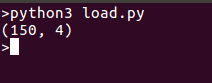### Scikit Learn SVM - Learning and Predicting

Now we have loaded data, let’s try learning from it and predict on new data. For this purpose we have to create an estimator and then call its fit method.

``````from sklearn import svm
from sklearn import datasets
clf = svm.LinearSVC()
# learn from the data
clf.fit(iris.data, iris.target)
# predict for unseen data
clf.predict([[ 5.0,  3.6,  1.3,  0.25]])
# Parameters of model can be changed by using the attributes ending with an underscore
print(clf.coef_ )
``````

Here is what we get when we run this script: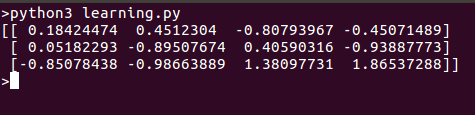### Scikit Learn Linear Regression

Creating various models is rather simple using scikit-learn. Let’s start with a simple example of regression.

``````#import the model
from sklearn import linear_model
reg = linear_model.LinearRegression()
# use it to fit a data
reg.fit ([[0, 0], [1, 1], [2, 2]], [0, 1, 2])
# Let's look into the fitted data
print(reg.coef_)
``````

Running the model should return a point that can be plotted on the same line: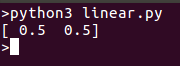### k-Nearest neighbour classifier

Let’s try a simple classification algorithm. This classifier uses an algorithm based on ball trees to represent the training samples.

``````from sklearn import datasets
# Create and fit a nearest-neighbor classifier
from sklearn import neighbors
knn = neighbors.KNeighborsClassifier()
knn.fit(iris.data, iris.target)
# Predict and print the result
result=knn.predict([[0.1, 0.2, 0.3, 0.4]])
print(result)
``````

Let’s run the classifier and check results, the classifier should return 0. Let’s try the example: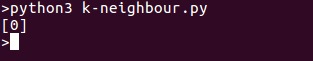### K-means clustering

This is the simplest clustering algorithm. The set is divided into ‘k’ clusters and each observation is assigned to a cluster. This is done iteratively until the clusters converge. We will create one such clustering model in the following program:

``````from sklearn import cluster, datasets
# create clusters for k=3
k=3
k_means = cluster.KMeans(k)
# fit data
k_means.fit(iris.data)
# print results
print( k_means.labels_[::10])
print( iris.target[::10])
``````

On running the program we’ll see separate clusters in the list. Here is the output for above code snippet: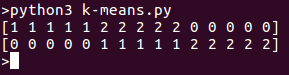## Conclusion

In this tutorial, we have seen that Scikit-Learn makes it easy to work with several machine learning algorithms. We have seen examples of Regression, Classification and Clustering. Scikit-Learn is still in development phase and being developed and maintained by volunteers but is very popular in community. Go and try your own examples.

]]>
Shubham 2022-08-03T18:17:40.812Z
<![CDATA[2 Easy Ways to Normalize data in Python]]> tag:www.digitalocean.com,2005:/community/tutorials/normalize-data-in-python 2022-08-03T17:28:27.417Z In this tutorial, we are going to learn about how to normalize data in Python. While normalizing we change the scale of the data. Data is most commonly rescaled to fall between 0-1.

## Why Do We Need To Normalize Data in Python?

Machine learning algorithms tend to perform better or converge faster when the different features (variables) are on a smaller scale. Therefore it is common practice to normalize the data before training machine learning models on it.

Normalization also makes the training process less sensitive to the scale of the features. This results in getting better coefficients after training.

This process of making features more suitable for training by rescaling is called feature scaling.

The formula for Normalization is given below :

We subtract the minimum value from each entry and then divide the result by the range. Where range is the difference between the maximum value and the minimum value.

## Steps to Normalize Data in Python

We are going to discuss two different ways to normalize data in python.

The first one is by using the method ‘normalize()’ under sklearn.

### Using normalize() from sklearn

Let’s start by importing processing from sklearn.

``````from sklearn import preprocessing
``````

Now, let’s create an array using Numpy.

``````import numpy as np
x_array = np.array([2,3,5,6,7,4,8,7,6])
``````

Now we can use the normalize() method on the array. This method normalizes data along a row. Let’s see the method in action.

``````normalized_arr = preprocessing.normalize([x_array])
print(normalized_arr)
``````

### Complete code

Here’s the complete code from this section :

``````from sklearn import preprocessing
import numpy as np
x_array = np.array([2,3,5,6,7,4,8,7,6])
normalized_arr = preprocessing.normalize([x_array])
print(normalized_arr)
``````

Output :

``````[0.11785113, 0.1767767 , 0.29462783, 0.35355339, 0.41247896,
0.23570226, 0.47140452, 0.41247896, 0.35355339]
``````

We can see that all the values are now between the range 0 to 1. This is how the normalize() method under sklearn works.

You can also normalize columns in a dataset using this method. Let’s see how to do that next.

### Normalize columns in a dataset using normalize()

Since normalize() only normalizes values along rows, we need to convert the column into an array before we apply the method.

To demonstrate we are going to use the California Housing dataset.

Let’s start by importing the dataset.

``````import pandas as pd
``````

Next, we need to pick a column and convert it into an array. We are going to use the 'total_bedrooms’ column.

``````from sklearn import preprocessing
x_array = np.array(housing['total_bedrooms'])
normalized_arr = preprocessing.normalize([x_array])
print(normalized_arr)
``````

Output :

``````[[0.01437454 0.02129852 0.00194947 ... 0.00594924 0.00618453 0.00336115]]
``````

### How to Normalize a Dataset Without Converting Columns to Array?

Let’s see what happens when we try to normalize a dataset without converting features into arrays for processing.

``````from sklearn import preprocessing
import pandas as pd
d = preprocessing.normalize(housing)
scaled_df = pd.DataFrame(d, columns=names)
``````

Output :

Here the values are normalized along the rows, which can be very unintuitive. Normalizing along rows means that each individual sample is normalized instead of the features.

However, you can specify the axis while calling the method to normalize along a feature (column).

The value of axis parameter is set to 1 by default. If we change the value to 0, the process of normalization happens along a column.

``````from sklearn import preprocessing
import pandas as pd
d = preprocessing.normalize(housing, axis=0)
scaled_df = pd.DataFrame(d, columns=names)
``````

Output :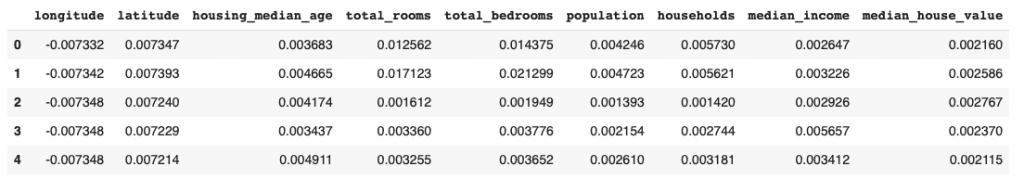You can see that the column for total_bedrooms in the output matches the one we got above after converting it into an array and then normalizing.

### Using MinMaxScaler() to Normalize Data in Python

Sklearn provides another option when it comes to normalizing data: MinMaxScaler.

This is a more popular choice for normalizing datasets.

Here’s the code for normalizing the housing dataset using MinMaxScaler :

``````from sklearn import preprocessing
import pandas as pd
scaler = preprocessing.MinMaxScaler()
names = housing.columns
d = scaler.fit_transform(housing)
scaled_df = pd.DataFrame(d, columns=names)
``````

Output :

You can see that the values in the output are between (0 and 1).

MinMaxScaler also gives you the option to select feature range. By default, the range is set to (0,1). Let’s see how to change the range to (0,2).

``````from sklearn import preprocessing
import pandas as pd
scaler = preprocessing.MinMaxScaler(feature_range=(0, 2))
names = housing.columns
d = scaler.fit_transform(housing)
scaled_df = pd.DataFrame(d, columns=names)
``````

Output :

The values in the output are now between (0,2).

## Conclusion

These are two methods to normalize data in Python. We covered two methods of normalizing data under sklearn. Hope you had fun learning with us!

]]>
Jayant Verma 2022-08-03T17:28:27.417Z
<![CDATA[An Introduction to Machine Learning]]> tag:www.digitalocean.com,2005:/community/tutorials/an-introduction-to-machine-learning 2022-05-31T18:26:30.305Z Introduction

Machine learning is a subfield of artificial intelligence (AI). The goal of machine learning generally is to understand the structure of data and fit that data into models that can be understood and utilized by people.

Although machine learning is a field within computer science, it differs from traditional computational approaches. In traditional computing, algorithms are sets of explicitly programmed instructions used by computers to calculate or problem solve. Machine learning algorithms instead allow for computers to train on data inputs and use statistical analysis in order to output values that fall within a specific range. Because of this, machine learning facilitates computers in building models from sample data in order to automate decision-making processes based on data inputs.

Any technology user today has benefitted from machine learning. Facial recognition technology allows social media platforms to help users tag and share photos of friends. Optical character recognition (OCR) technology converts images of text into movable type. Recommendation engines, powered by machine learning, suggest what movies or television shows to watch next based on user preferences. Self-driving cars that rely on machine learning to navigate may soon be available to consumers.

Machine learning is a continuously developing field. Because of this, there are some considerations to keep in mind as you work with machine learning methodologies, or analyze the impact of machine learning processes.

In this tutorial, we’ll look into the common machine learning methods of supervised and unsupervised learning, and common algorithmic approaches in machine learning, including the k-nearest neighbor algorithm, decision tree learning, and deep learning. We’ll explore which programming languages are most used in machine learning, providing you with some of the positive and negative attributes of each. Additionally, we’ll discuss biases that are perpetuated by machine learning algorithms, and consider what can be kept in mind to prevent these biases when building algorithms.

## Machine Learning Methods

In machine learning, tasks are generally classified into broad categories. These categories are based on how learning is received or how feedback on the learning is given to the system developed.

Two of the most widely adopted machine learning methods are supervised learning which trains algorithms based on example input and output data that is labeled by humans, and unsupervised learning which provides the algorithm with no labeled data in order to allow it to find structure within its input data. Let’s explore these methods in more detail.

### Supervised Learning

In supervised learning, the computer is provided with example inputs that are labeled with their desired outputs. The purpose of this method is for the algorithm to be able to “learn” by comparing its actual output with the “taught” outputs to find errors, and modify the model accordingly. Supervised learning therefore uses patterns to predict label values on additional unlabeled data.

For example, with supervised learning, an algorithm may be fed data with images of sharks labeled as `fish` and images of oceans labeled as `water`. By being trained on this data, the supervised learning algorithm should be able to later identify unlabeled shark images as `fish` and unlabeled ocean images as `water`.

A common use case of supervised learning is to use historical data to predict statistically likely future events. It may use historical stock market information to anticipate upcoming fluctuations, or be employed to filter out spam emails. In supervised learning, tagged photos of dogs can be used as input data to classify untagged photos of dogs.

### Unsupervised Learning

In unsupervised learning, data is unlabeled, so the learning algorithm is left to find commonalities among its input data. As unlabeled data are more abundant than labeled data, machine learning methods that facilitate unsupervised learning are particularly valuable.

The goal of unsupervised learning may be as straightforward as discovering hidden patterns within a dataset, but it may also have a goal of feature learning, which allows the computational machine to automatically discover the representations that are needed to classify raw data.

Unsupervised learning is commonly used for transactional data. You may have a large dataset of customers and their purchases, but as a human you will likely not be able to make sense of what similar attributes can be drawn from customer profiles and their types of purchases. With this data fed into an unsupervised learning algorithm, it may be determined that women of a certain age range who buy unscented soaps are likely to be pregnant, and therefore a marketing campaign related to pregnancy and baby products can be targeted to this audience in order to increase their number of purchases.

Without being told a “correct” answer, unsupervised learning methods can look at complex data that is more expansive and seemingly unrelated in order to organize it in potentially meaningful ways. Unsupervised learning is often used for anomaly detection including for fraudulent credit card purchases, and recommender systems that recommend what products to buy next. In unsupervised learning, untagged photos of dogs can be used as input data for the algorithm to find likenesses and classify dog photos together.

## Approaches

As a field, machine learning is closely related to computational statistics, so having a background knowledge in statistics is useful for understanding and leveraging machine learning algorithms.

For those who may not have studied statistics, it can be helpful to first define correlation and regression, as they are commonly used techniques for investigating the relationship among quantitative variables. Correlation is a measure of association between two variables that are not designated as either dependent or independent. Regression at a basic level is used to examine the relationship between one dependent and one independent variable. Because regression statistics can be used to anticipate the dependent variable when the independent variable is known, regression enables prediction capabilities.

Approaches to machine learning are continuously being developed. For our purposes, we’ll go through a few of the popular approaches that are being used in machine learning at the time of writing.

### k-nearest neighbor

The k-nearest neighbor algorithm is a pattern recognition model that can be used for classification as well as regression. Often abbreviated as k-NN, the k in k-nearest neighbor is a positive integer, which is typically small. In either classification or regression, the input will consist of the k closest training examples within a space.

We will focus on k-NN classification. In this method, the output is class membership. This will assign a new object to the class most common among its k nearest neighbors. In the case of k = 1, the object is assigned to the class of the single nearest neighbor.

Let’s look at an example of k-nearest neighbor. In the diagram below, there are blue diamond objects and orange star objects. These belong to two separate classes: the diamond class and the star class.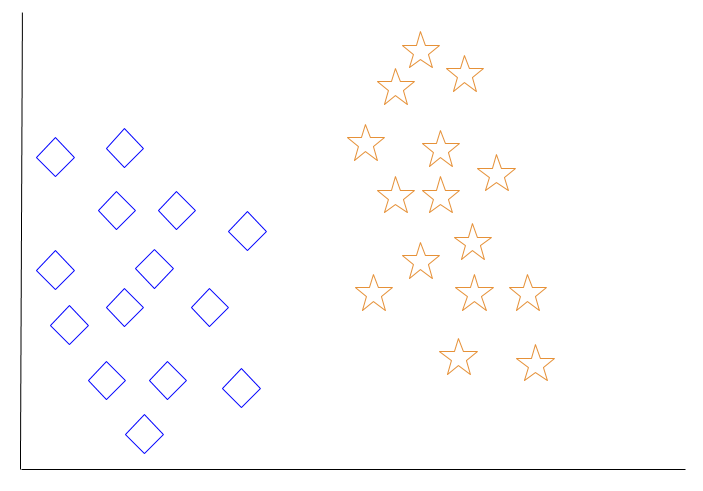When a new object is added to the space — in this case a green heart — we will want the machine learning algorithm to classify the heart to a certain class.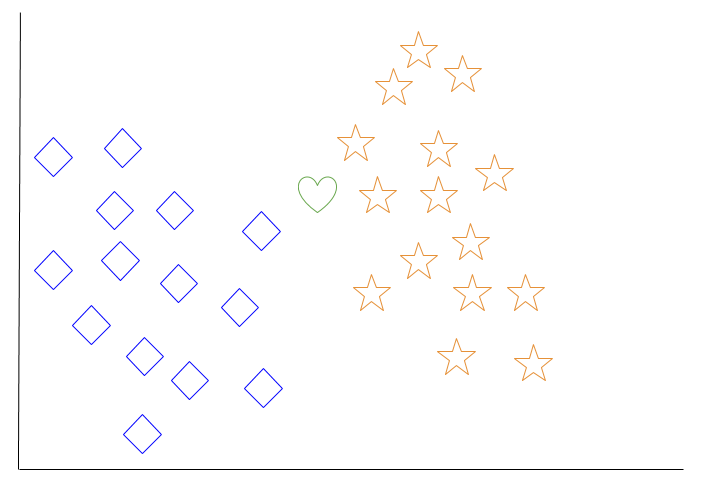When we choose k = 3, the algorithm will find the three nearest neighbors of the green heart in order to classify it to either the diamond class or the star class.

In our diagram, the three nearest neighbors of the green heart are one diamond and two stars. Therefore, the algorithm will classify the heart with the star class.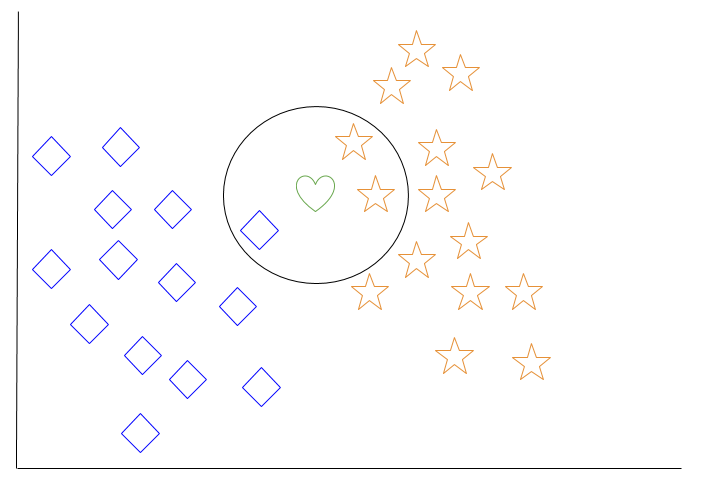Among the most basic of machine learning algorithms, k-nearest neighbor is considered to be a type of “lazy learning” as generalization beyond the training data does not occur until a query is made to the system.

### Decision Tree Learning

For general use, decision trees are employed to visually represent decisions and show or inform decision making. When working with machine learning and data mining, decision trees are used as a predictive model. These models map observations about data to conclusions about the data’s target value.

The goal of decision tree learning is to create a model that will predict the value of a target based on input variables.

In the predictive model, the data’s attributes that are determined through observation are represented by the branches, while the conclusions about the data’s target value are represented in the leaves.

When “learning” a tree, the source data is divided into subsets based on an attribute value test, which is repeated on each of the derived subsets recursively. Once the subset at a node has the equivalent value as its target value has, the recursion process will be complete.

Let’s look at an example of various conditions that can determine whether or not someone should go fishing. This includes weather conditions as well as barometric pressure conditions.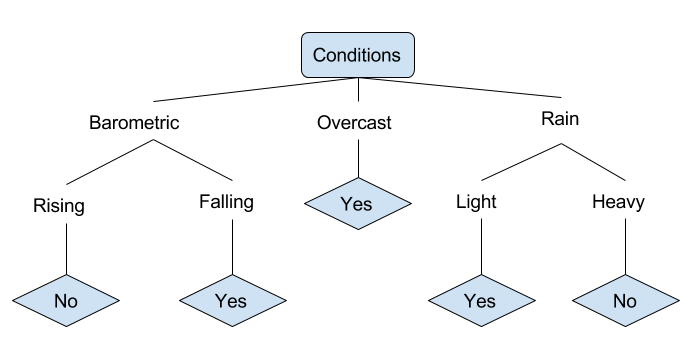In the simplified decision tree above, an example is classified by sorting it through the tree to the appropriate leaf node. This then returns the classification associated with the particular leaf, which in this case is either a `Yes` or a `No`. The tree classifies a day’s conditions based on whether or not it is suitable for going fishing.

A true classification tree data set would have a lot more features than what is outlined above, but relationships should be straightforward to determine. When working with decision tree learning, several determinations need to be made, including what features to choose, what conditions to use for splitting, and understanding when the decision tree has reached a clear ending.

### Deep Learning

Deep learning attempts to imitate how the human brain can process light and sound stimuli into vision and hearing. A deep learning architecture is inspired by biological neural networks and consists of multiple layers in an artificial neural network made up of hardware and GPUs.

Deep learning uses a cascade of nonlinear processing unit layers in order to extract or transform features (or representations) of the data. The output of one layer serves as the input of the successive layer. In deep learning, algorithms can be either supervised and serve to classify data, or unsupervised and perform pattern analysis.

Among the machine learning algorithms that are currently being used and developed, deep learning absorbs the most data and has been able to beat humans in some cognitive tasks. Because of these attributes, deep learning has become an approach with significant potential in the artificial intelligence space

Computer vision and speech recognition have both realized significant advances from deep learning approaches. IBM Watson is a well-known example of a system that leverages deep learning.

## Programming Languages

When choosing a language to specialize in with machine learning, you may want to consider the skills listed on current job advertisements as well as libraries available in various languages that can be used for machine learning processes.

Python’s is one of the most popular languages for working with machine learning due to the many available frameworks, including TensorFlow, PyTorch, and Keras. As a language that has readable syntax and the ability to be used as a scripting language, Python proves to be powerful and straightforward both for preprocessing data and working with data directly. The scikit-learn machine learning library is built on top of several existing Python packages that Python developers may already be familiar with, namely NumPy, SciPy, and Matplotlib.

To get started with Python, you can read our tutorial series on “How To Code in Python 3,” or read specifically on “How To Build a Machine Learning Classifier in Python with scikit-learn” or “How To Perform Neural Style Transfer with Python 3 and PyTorch.”

Java is widely used in enterprise programming, and is generally used by front-end desktop application developers who are also working on machine learning at the enterprise level. Usually it is not the first choice for those new to programming who want to learn about machine learning, but is favored by those with a background in Java development to apply to machine learning. In terms of machine learning applications in industry, Java tends to be used more than Python for network security, including in cyber attack and fraud detection use cases.

Among machine learning libraries for Java are Deeplearning4j, an open-source and distributed deep-learning library written for both Java and Scala; MALLET (MAchine Learning for LanguagE Toolkit) allows for machine learning applications on text, including natural language processing, topic modeling, document classification, and clustering; and Weka, a collection of machine learning algorithms to use for data mining tasks.

C++ is the language of choice for machine learning and artificial intelligence in game or robot applications (including robot locomotion). Embedded computing hardware developers and electronics engineers are more likely to favor C++ or C in machine learning applications due to their proficiency and level of control in the language. Some machine learning libraries you can use with C++ include the scalable mlpack, Dlib offering wide-ranging machine learning algorithms, and the modular and open-source Shark.

## Human Biases

Although data and computational analysis may make us think that we are receiving objective information, this is not the case; being based on data does not mean that machine learning outputs are neutral. Human bias plays a role in how data is collected, organized, and ultimately in the algorithms that determine how machine learning will interact with that data.

If, for example, people are providing images for “fish” as data to train an algorithm, and these people overwhelmingly select images of goldfish, a computer may not classify a shark as a fish. This would create a bias against sharks as fish, and sharks would not be counted as fish.

When using historical photographs of scientists as training data, a computer may not properly classify scientists who are also people of color or women. In fact, recent peer-reviewed research has indicated that AI and machine learning programs exhibit human-like biases that include race and gender prejudices. See, for example “Semantics derived automatically from language corpora contain human-like biases” and “Men Also Like Shopping: Reducing Gender Bias Amplification using Corpus-level Constraints” [PDF].

As machine learning is increasingly leveraged in business, uncaught biases can perpetuate systemic issues that may prevent people from qualifying for loans, from being shown ads for high-paying job opportunities, or from receiving same-day delivery options.

Because human bias can negatively impact others, it is extremely important to be aware of it, and to also work towards eliminating it as much as possible. One way to work towards achieving this is by ensuring that there are diverse people working on a project and that diverse people are testing and reviewing it. Others have called for regulatory third parties to monitor and audit algorithms, building alternative systems that can detect biases, and ethics reviews as part of data science project planning. Raising awareness about biases, being mindful of our own unconscious biases, and structuring equity in our machine learning projects and pipelines can work to combat bias in this field.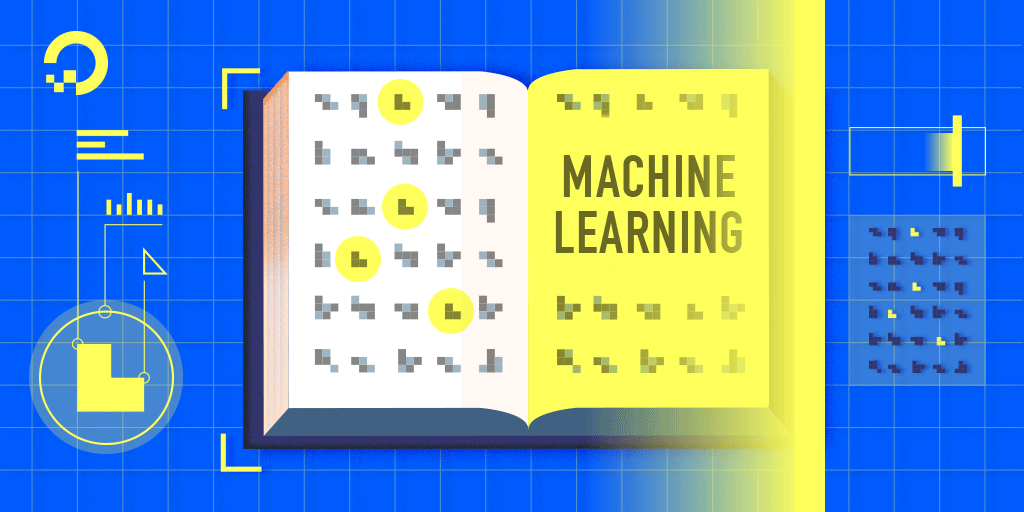## Conclusion

This tutorial reviewed some of the use cases of machine learning, common methods and popular approaches used in the field, suitable machine learning programming languages, and also covered some things to keep in mind in terms of unconscious biases being replicated in algorithms.

Because machine learning is a field that is continuously being innovated, it is important to keep in mind that algorithms, methods, and approaches will continue to change.

In addition to reading our tutorials on “How To Build a Machine Learning Classifier in Python with scikit-learn” or “How To Perform Neural Style Transfer with Python 3 and PyTorch,” you can learn more about working with data in the technology industry by reading our Data Analysis tutorials.

]]>
Lisa Tagliaferri 2022-05-31T18:26:30.305Z
<![CDATA[A question related to the tutorial of How To Build a Deep Learning Model to Predict Employee Retention Using Keras and TensorFlow]]> tag:www.digitalocean.com,2005:/community/questions/a-question-related-to-the-tutorial-of-how-to-build-a-deep-learning-model-to-predict-employee-retention-using-keras-and-tensorflow 2022-05-05T10:43:17.277Z Firstly thanks for the tutorial and explanation of How To Build a Deep Learning Model to Predict Employee Retention Using Keras and TensorFlow. This is more related to the coding part which i was trying from the tutorial .At the step of hyperparameter tuning, when fiting the model , grid_search = grid_search.fit(X_train,y_train) , its actually throwing the error as TypeError: make_classifier() missing 1 required positional argument: ‘optimizer’ Could you please guide on this error

]]>
HappyDarkBlueWalrus 2022-05-05T10:43:17.277Z
<![CDATA[How To Install the Anaconda Python Distribution on Ubuntu 22.04]]> tag:www.digitalocean.com,2005:/community/tutorials/how-to-install-the-anaconda-python-distribution-on-ubuntu-22-04 2022-04-26T14:40:27.830Z Introduction

Anaconda is an open-source package manager, environment manager, and distribution of the Python and R programming languages. It is commonly used for data science, machine learning, large-scale data processing, scientific computing, and predictive analytics.

Offering a collection of over 1,000 data science packages, Anaconda is available in both free and paid enterprise versions. The Anaconda distribution ships with the `conda` command-line utility. You can learn more about Anaconda and `conda` by reading the official Anaconda Documentation.

This tutorial will guide you through installing the Python 3 version of Anaconda on an Ubuntu 22.04 server.

## Prerequisites

Before you begin with this guide, you should have:

## Installing Anaconda

The best way to install Anaconda is to download the latest Anaconda installer bash script, verify it, and then run it.

Find the latest version of Anaconda for Python 3 at the Anaconda Downloads page. At the time of writing, the latest version is 2021.11, but you should use a later stable version if it is available.

Next, change to the `/tmp` directory on your server. This is a good directory to download ephemeral items, like the Anaconda bash script, which you won’t need after running it.

``````cd /tmp

``````

Use `curl` to download the link that you copied from the Anaconda website. You’ll output this to a file called `anaconda.sh` for quicker use.

``````curl https://repo.anaconda.com/archive/Anaconda3-2021.11-Linux-x86_64.sh --output anaconda.sh

``````

You can now verify the data integrity of the installer with cryptographic hash verification through the SHA-256 checksum. You’ll use the `sha256sum` command along with the filename of the script:

``````sha256sum anaconda.sh

``````

You’ll receive output that looks similar to this:

``````Outputfedf9e340039557f7b5e8a8a86affa9d299f5e9820144bd7b92ae9f7ee08ac60  anaconda.sh
``````

You should check the output against the hashes available at the Anaconda with Python 3 on 64-bit Linux page for your appropriate Anaconda version. As long as your output matches the hash displayed in the `sha256` row, you’re good to go.

Now you can run the script:

``````bash anaconda.sh

``````

``````Output
Welcome to Anaconda3 2021.11

agreement.
>>>
``````

Press `ENTER` to continue and then press `ENTER` to read through the license. Once you’re done reading the license, you’ll be prompted to approve the license terms:

``````OutputDo you approve the license terms? [yes|no]
``````

As long as you agree, type `yes`.

At this point, you’ll be prompted to choose the location of the installation. You can press `ENTER` to accept the default location, or specify a different location to modify it.

``````OutputAnaconda3 will now be installed into this location:
/home/sammy/anaconda3

- Press ENTER to confirm the location
- Press CTRL-C to abort the installation
- Or specify a different location below

[/home/sammy/anaconda3] >>>
``````

The installation process will continue. Note that it may take some time.

Once installation is complete, you’ll receive the following output:

``````Output...
installation finished.
Do you wish the installer to initialize Anaconda3
by running conda init? [yes|no]
[no] >>>
``````

Type `yes` so that you can initialize Anaconda3. You’ll receive some output that states changes made in various directories. One of the lines you receive will thank you for installing Anaconda.

``````Output...
Thank you for installing Anaconda3!
...
``````

You can now activate the installation by sourcing the `~/.bashrc` file:

``````source ~/.bashrc

``````

Once you have done that, you’ll be placed into the default `base` programming environment of Anaconda, and your command prompt will change to be the following:

``````

``````

Although Anaconda ships with this default `base` programming environment, you should create separate environments for your programs and to keep them isolated from each other.

You can further verify your install by making use of the `conda` command, for example with `list`:

``````conda list

``````

You’ll receive output of all the packages you have available through the Anaconda installation:

``````Output# packages in environment at /home/sammy/anaconda3:
#
# Name                    Version                   Build  Channel
_ipyw_jlab_nb_ext_conf    0.1.0            py39h06a4308_0
_libgcc_mutex             0.1                        main
_openmp_mutex             4.5                       1_gnu
alabaster                 0.7.12             pyhd3eb1b0_0
anaconda                  2021.11                  py39_0
...
``````

Now that Anaconda is installed, you can go on to setting up Anaconda environments.

## Setting Up Anaconda Environments

Anaconda virtual environments allow you to keep projects organized by Python versions and packages needed. For each Anaconda environment you set up, you can specify which version of Python to use and can keep all of your related programming files together within that directory.

First, you can check to see which versions of Python are available for us to use:

``````conda search "^python\$"

``````

You’ll receive output with the different versions of Python that you can target, including both Python 3 and Python 2 versions. Since you are using the Anaconda with Python 3 in this tutorial, you will have access only to the Python 3 versions of packages.

Next, create an environment using the most recent version of Python 3. You can achieve this by assigning version 3 to the `python` argument. You’ll call the environment my_env, but you’ll likely want to use a more descriptive name for your environment especially if you are using environments to access more than one version of Python.

``````conda create --name my_env python=3

``````

You’ll receive output with information about what is downloaded and which packages will be installed, and then be prompted to proceed with `y` or `n`. As long as you agree, type `y`.

The `conda` utility will now fetch the packages for the environment and let you know when it’s complete.

You can activate your new environment by typing the following:

``````conda activate my_env

``````

With your environment activated, your command prompt prefix will reflect that you are no longer in the `base` environment, but in the new one that you just created.

``````

``````

Within the environment, you can verify that you’re using the version of Python that you had intended to use:

`````` python --version

``````
``````OutputPython 3.10.4
``````

When you’re ready to deactivate your Anaconda environment, you can do so by typing:

``````conda deactivate

``````

Note that you can replace the word `source` with `.` to achieve the same results.

To target a more specific version of Python, you can pass a specific version to the `python` argument, like `3.5`, for example:

``````conda create -n my_env35 python=3.5

``````

You can inspect all of the environments you have set up with this command:

``````conda info --envs

``````
``````Output# conda environments:
#
base                  *  /home/sammy/anaconda3
my_env                   /home/sammy/anaconda3/envs/my_env
my_env35                 /home/sammy/anaconda3/envs/my_env35

``````

The asterisk indicates the current active environment.

Each environment you create with `conda create` will come with several default packages:

• `_libgcc_mutex`
• `ca-certificates`
• `certifi`
• `libedit`
• `libffi`
• `libgcc-ng`
• `libstdcxx-ng`
• `ncurses`
• `openssl`
• `pip`
• `python`
• `readline`
• `setuptools`
• `sqlite`
• `tk`
• `wheel`
• `xz`
• `zlib`

You can add additional packages, such as `numpy` for example, with the following command:

``````conda install --name my_env35 numpy

``````

If you know you would like a `numpy` environment upon creation, you can target it in your `conda create` command:

``````conda create --name my_env python=3 numpy

``````

If you are no longer working on a specific project and have no further need for the associated environment, you can remove it. To do so, type the following:

``````conda remove --name my_env35 --all

``````

Now, when you type the `conda info --envs` command, the environment that you removed will no longer be listed.

## Updating Anaconda

You should regularly ensure that Anaconda is up-to-date so that you are working with all the latest package releases.

To do this, you should first update the `conda` utility:

``````conda update conda

``````

When prompted to do so, type `y` to proceed with the update.

Once the update of `conda` is complete, you can update the Anaconda distribution:

``````conda update anaconda

``````

Again, when prompted to do so, type `y` to proceed.

This will ensure that you are using the latest releases of `conda` and Anaconda.

## Uninstalling Anaconda

If you are no longer using Anaconda and find that you need to uninstall it, you should start with the `anaconda-clean` module, which will remove configuration files for when you uninstall Anaconda.

``````conda install anaconda-clean

``````

Type `y` when prompted to do so.

Once it is installed, you can run the following command. You will be prompted to answer `y` before deleting each one. If you would prefer not to be prompted, add `--yes` to the end of your command:

``````anaconda-clean

``````

This will also create a backup folder called `.anaconda_backup` in your home directory:

``````OutputBackup directory: /home/sammy/.anaconda_backup/2022-03-31T182409
``````

You can now remove your entire Anaconda directory by entering the following command:

``````rm -rf ~/anaconda3

``````

Finally, you can remove the PATH line from your `.bashrc` file that Anaconda added. To do so, first open a text editor such as nano:

``````nano ~/.bashrc

``````

Then scroll down to the end of the file (if this is a recent install) or type `CTRL + W` to search for Anaconda. Delete or comment out this Anaconda block:

/home/sammy/.bashrc
``````...
# >>> conda initialize >>>
# !! Contents within this block are managed by 'conda init' !!
__conda_setup="\$('/home/sammy/anaconda3/bin/conda' 'shell.bash' 'hook' 2> /dev/null)"
if [ \$? -eq 0 ]; then
eval "\$__conda_setup"
else
if [ -f "/home/sammy/anaconda3/etc/profile.d/conda.sh" ]; then
. "/home/sammy/anaconda3/etc/profile.d/conda.sh"
else
export PATH="/home/sammy/anaconda3/bin:\$PATH"
fi
fi
unset __conda_setup
# <<< conda initialize <<<
``````

When you’re done editing the file, type `CTRL + X` to exit and `y` to save changes.

Anaconda is now removed from your server. If you did not deactivate the `base` programming environment, you can exit and re-enter the server to remove it.

## Conclusion

You have completed the installation of Anaconda, working with the `conda` command-line utility, setting up environments, updating Anaconda, and deleting Anaconda if you no longer need it.

You can use Anaconda to help you manage workloads for data science, scientific computing, analytics, and large-scale data processing. From here, you can check out tutorials on data analysis and machine learning to learn more about various tools available to use and projects that you can do.

We also have a free machine learning ebook available for download, Python Machine Learning Projects.

]]>
Lisa Tagliaferri Tony Tran 2022-04-26T14:40:27.830Z
<![CDATA[How To Build a Deep Learning Model to Predict Employee Retention Using Keras and TensorFlow]]> tag:www.digitalocean.com,2005:/community/tutorials/how-to-build-a-deep-learning-model-to-predict-employee-retention-using-keras-and-tensorflow 2022-04-04T08:55:00.268Z The author selected Girls Who Code to receive a donation as part of the Write for DOnations program.

### Introduction

Keras is a neural network API that is written in Python. It runs on top of TensorFlow, CNTK, or Theano. It is a high-level abstraction of these deep learning frameworks and therefore makes experimentation faster and easier. Keras is modular, which means implementation is seamless as developers can quickly extend models by adding modules.

TensorFlow is an open-source software library for machine learning. It works efficiently with computation involving arrays; so it’s a great choice for the model you’ll build in this tutorial. Furthermore, TensorFlow allows for the execution of code on either CPU or GPU, which is a useful feature especially when you’re working with a massive dataset.

In this tutorial, you’ll build a deep learning model that will predict the probability of an employee leaving a company. Retaining the best employees is an important factor for most organizations. To build your model, you’ll use this dataset available at Kaggle, which has features that measure employee satisfaction in a company. To create this model, you’ll use the Keras sequential layer to build the different layers for the model.

## Prerequisites

Before you begin this tutorial you’ll need the following:

## Step 1 — Data Pre-processing

Data Pre-processing is necessary to prepare your data in a manner that a deep learning model can accept. If there are categorical variables in your data, you have to convert them to numbers because the algorithm only accepts numerical figures. A categorical variable represents quantitive data represented by names. In this step, you’ll load in your dataset using `pandas`, which is a data manipulation Python library.

Before you begin data pre-processing, you’ll activate your environment and ensure you have all the necessary packages installed to your machine. It’s advantageous to use `conda` to install `keras` and `tensorflow` since it will handle the installation of any necessary dependencies for these packages, and ensure they are compatible with `keras` and `tensorflow`. In this way, using the Anaconda Python distribution is a good choice for data science related projects.

Move into the environment you created in the prerequisite tutorial:

``````conda activate my_env

``````

Run the following command to install `keras` and `tensorflow`:

``````conda install tensorflow keras

``````

Now, open Jupyter Notebook to get started. Jupyter Notebook is opened by typing the following command on your terminal:

``````jupyter notebook

``````

Note: If you’re working from a remote server, you’ll need to use SSH tunneling to access your notebook. Please revisit step 2 of the prerequisite tutorial for detailed on instructions on setting up SSH tunneling. You can use the following command from your local machine to initiate your SSH tunnel:

``````ssh -L 8888:localhost:8888 your_username@your_server_ip

``````

After accessing Jupyter Notebook, click on the anaconda3 file, and then click New at the top of the screen, and select Python 3 to load a new notebook.

Now, you’ll import the required modules for the project and then load the dataset in a notebook cell. You’ll load in the `pandas` module for manipulating your data and `numpy` for converting the data into `numpy` arrays. You’ll also convert all the columns that are in string format to numerical values for your computer to process.

Insert the following code into a notebook cell and then click Run:

``````import pandas as pd
import numpy as np
``````

You’ve imported `numpy` and `pandas`. You then used `pandas` to load in the dataset for the model.

You can get a glimpse at the dataset you’re working with by using `head()`. This is a useful function from `pandas` that allows you to view the first five records of your dataframe. Add the following code to a notebook cell and then run it:

``````df.head()
``````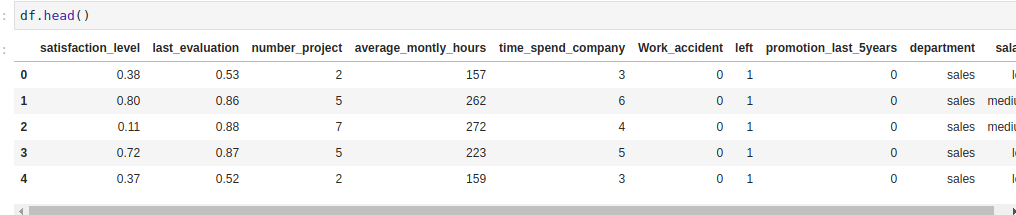You’ll now proceed to convert the categorical columns to numbers. You do this by converting them to dummy variables. Dummy variables are usually ones and zeros that indicate the presence or absence of a categorical feature. In this kind of situation, you also avoid the dummy variable trap by dropping the first dummy.

Note: The dummy variable trap is a situation whereby two or more variables are highly correlated. This leads to your model performing poorly. You, therefore, drop one dummy variable to always remain with N-1 dummy variables. Any of the dummy variables can be dropped because there is no preference as long as you remain with N-1 dummy variables. An example of this is if you were to have an on/off switch. When you create the dummy variable you shall get two columns: an `on` column and an `off` column. You can drop one of the columns because if the switch isn’t on, then it is off.

Insert this code in the next notebook cell and execute it:

``````feats = ['department','salary']
df_final = pd.get_dummies(df,columns=feats,drop_first=True)
``````

`feats = ['department','salary']` defines the two columns for which you want to create dummy variables. `pd.get_dummies(df,columns=feats,drop_first=True)` will generate the numerical variables that your employee retention model requires. It does this by converting the `feats` that you define from categorical to numerical variables.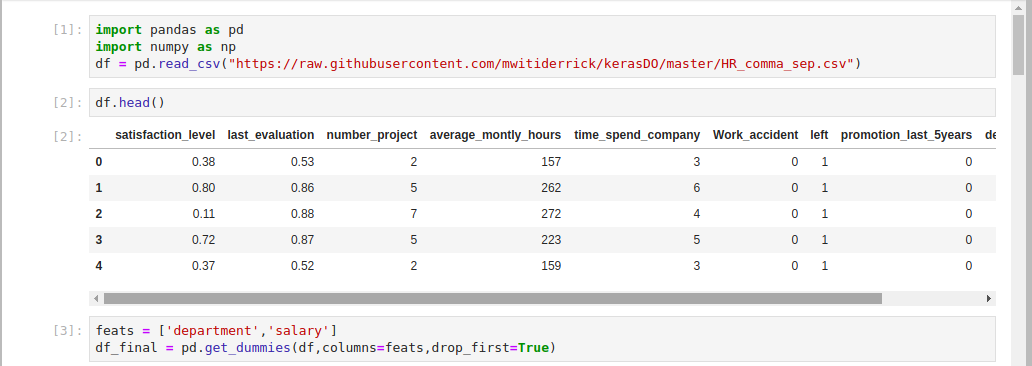You’ve loaded in the dataset and converted the salary and department columns into a format the `keras` deep learning model can accept. In the next step, you will split the dataset into a training and testing set.

## Step 2 — Separating Your Training and Testing Datasets

You’ll use `scikit-learn` to split your dataset into a training and a testing set. This is necessary so you can use part of the employee data to train the model and a part of it to test its performance. Splitting a dataset in this way is a common practice when building deep learning models.

It is important to implement this split in the dataset so the model you build doesn’t have access to the testing data during the training process. This ensures that the model learns only from the training data, and you can then test its performance with the testing data. If you exposed your model to testing data during the training process then it would memorize the expected outcomes. Consequently, it would fail to give accurate predictions on data that it hasn’t seen.

You’ll start by importing the `train_test_split` module from the `scikit-learn` package. This is the module that will provide the splitting functionality. Insert this code in the next notebook cell and run:

``````from sklearn.model_selection import train_test_split
``````

With the `train_test_split` module imported, you’ll use the `left` column in your dataset to predict if an employee will leave the company. Therefore, it is essential that your deep learning model doesn’t come into contact with this column. Insert the following into a cell to drop the `left` column:

``````X = df_final.drop(['left'],axis=1).values
y = df_final['left'].values
``````

Your deep learning model expects to get the data as arrays. Therefore you use `numpy` to convert the data to `numpy` arrays with the `.values` attribute.

You’re now ready to convert the dataset into a testing and training set. You’ll use 70% of the data for training and 30% for testing. The training ratio is more than the testing ratio because you’ll need to use most of the data for the training process. If desired, you can also experiment with a ratio of 80% for the training set and 20% for the testing set.

Now add this code to the next cell and run to split your training and testing data to the specified ratio:

``````X_train, X_test, y_train, y_test = train_test_split(X, y, test_size=0.3)
``````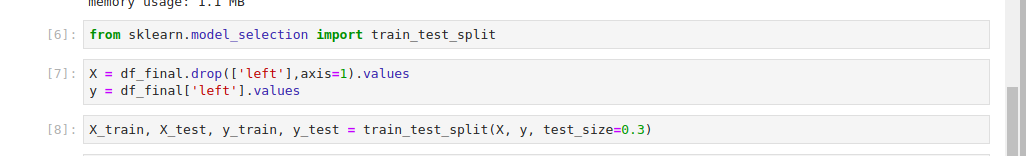You have now converted the data into the type that Keras expects it to be in (`numpy` arrays), and your data is split into a training and testing set. You’ll pass this data to the `keras` model later in the tutorial. Beforehand you need to transform the data, which you’ll complete in the next step.

## Step 3 — Transforming the Data

When building deep learning models it is usually good practice to scale your dataset in order to make the computations more efficient. In this step, you’ll scale the data using the `StandardScaler`; this will ensure that your dataset values have a mean of zero and a unit variable. This transforms the dataset to be normally distributed. You’ll use the `scikit-learn` `StandardScaler` to scale the features to be within the same range. This will transform the values to have a mean of 0 and a standard deviation of 1. This step is important because you’re comparing features that have different measurements; so it is typically required in machine learning.

To scale the training set and the test set, add this code to the notebook cell and run it:

``````from sklearn.preprocessing import StandardScaler
sc = StandardScaler()
X_train = sc.fit_transform(X_train)
X_test = sc.transform(X_test)
``````

Here, you start by importing the `StandardScaler` and calling an instance of it. You then use its `fit_transform` method to scale the training and testing set.

You have scaled all your dataset features to be within the same range. You can start building the artificial neural network in the next step.

## Step 4 — Building the Artificial Neural Network

Now you will use `keras` to build the deep learning model. To do this, you’ll import `keras`, which will use `tensorflow` as the backend by default. From `keras`, you’ll then import the `Sequential` module to initialize the artificial neural network. An artificial neural network is a computational model that is built using inspiration from the workings of the human brain. You’ll import the `Dense` module as well, which will add layers to your deep learning model.

When building a deep learning model you usually specify three layer types:

• The input layer is the layer to which you’ll pass the features of your dataset. There is no computation that occurs in this layer. It serves to pass features to the hidden layers.
• The hidden layers are usually the layers between the input layer and the output layer—and there can be more than one. These layers perform the computations and pass the information to the output layer.
• The output layer represents the layer of your neural network that will give you the results after training your model. It is responsible for producing the output variables.

To import the `Keras`, `Sequential`, and `Dense` modules, run the following code in your notebook cell:

``````import keras
from keras.models import Sequential
from keras.layers import Dense
``````

You’ll use `Sequential` to initialize a linear stack of layers. Since this is a classification problem, you’ll create a classifier variable. A classification problem is a task where you have labeled data and would like to make some predictions based on the labeled data. Add this code to your notebook to create a classifier variable:

``````classifier = Sequential()
``````

You’ve used `Sequential` to initialize the classifier.

``````classifier.add(Dense(9, kernel_initializer = "uniform",activation = "relu", input_dim=18))
``````

You add layers using the `.add()` function on your classifier and specify some parameters:

• The first parameter is the number of nodes that your network should have. The connection between different nodes is what forms the neural network. One of the strategies to determine the number of nodes is to take the average of the nodes in the input layer and the output layer.

• The second parameter is the `kernel_initializer.` When you fit your deep learning model the weights will be initialized to numbers close to zero, but not zero. To achieve this you use the uniform distribution initializer. `kernel_initializer` is the function that initializes the weights.

• The third parameter is the `activation` function. Your deep learning model will learn through this function. There are usually linear and non-linear activation functions. You use the `relu` activation function because it generalizes well on your data. Linear functions are not good for problems like these because they form a straight line.

• The last parameter is `input_dim`, which represents the number of features in your dataset.

Now you’ll add the output layer that will give you the predictions:

``````classifier.add(Dense(1, kernel_initializer = "uniform",activation = "sigmoid"))
``````

The output layer takes the following parameters:

• The number of output nodes. You expect to get one output: if an employee leaves the company. Therefore you specify one output node.

• For `kernel_initializer` you use the `sigmoid` activation function so that you can get the probability that an employee will leave. In the event that you were dealing with more than two categories, you would use the `softmax` activation function, which is a variant of the `sigmoid` activation function.

Next, you’ll apply a gradient descent to the neural network. This is an optimization strategy that works to reduce errors during the training process. Gradient descent is how randomly assigned weights in a neural network are adjusted by reducing the cost function, which is a measure of how well a neural network performs based on the output expected from it.

The aim of a gradient descent is to get the point where the error is at its least. This is done by finding where the cost function is at its minimum, which is referred to as a local minimum. In gradient descent, you differentiate to find the slope at a specific point and find out if the slope is negative or positive—you’re descending into the minimum of the cost function. There are several types of optimization strategies, but you’ll use a popular one known as `adam` in this tutorial.

``````classifier.compile(optimizer= "adam",loss = "binary_crossentropy",metrics = ["accuracy"])
``````

Applying gradient descent is done via the `compile` function that takes the following parameters:

• `optimizer` is the gradient descent.
• `loss` is a function that you’ll use in the gradient descent. Since this is a binary classification problem you use the `binary_crossentropy` `loss` function.
• The last parameter is the `metric` that you’ll use to evaluate your model. In this case, you’d like to evaluate it based on its accuracy when making predictions.

You’re ready to fit your classifier to your dataset. Keras makes this possible via the `.fit()` method. To do this, insert the following code into your notebook and run it in order to fit the model to your dataset:

``````classifier.fit(X_train, y_train, batch_size = 10, epochs = 1)
``````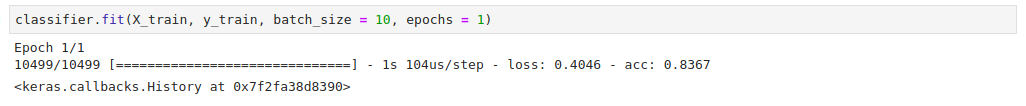The `.fit()` method takes a couple of parameters:

• The first parameter is the training set with the features.

• The second parameter is the column that you’re making the predictions on.

• The `batch_size` represents the number of samples that will go through the neural network at each training round.

• `epochs` represents the number of times that the dataset will be passed via the neural network. The more epochs the longer it will take to run your model, which also gives you better results.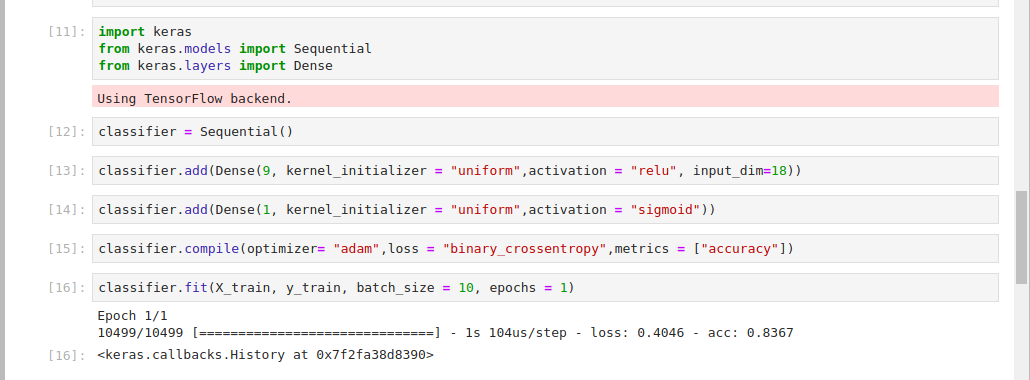You’ve created your deep learning model, compiled it, and fitted it to your dataset. You’re ready to make some predictions using the deep learning model. In the next step, you’ll start making predictions with the dataset that the model hasn’t yet seen.

## Step 5 — Running Predictions on the Test Set

To start making predictions, you’ll use the testing dataset in the model that you’ve created. Keras enables you to make predictions by using the `.predict()` function.

Insert the following code in the next notebook cell to begin making predictions:

``````y_pred = classifier.predict(X_test)
``````

Since you’ve already trained the classifier with the training set, this code will use the learning from the training process to make predictions on the test set. This will give you the probabilities of an employee leaving. You’ll work with a probability of 50% and above to indicate a high chance of the employee leaving the company.

Enter the following line of code in your notebook cell in order to set this threshold:

``````y_pred = (y_pred > 0.5)
``````

You’ve created predictions using the predict method and set the threshold for determining if an employee is likely to leave. To evaluate how well the model performed on the predictions, you will next use a confusion matrix.

## Step 6 — Checking the Confusion Matrix

In this step, you will use a confusion matrix to check the number of correct and incorrect predictions. A confusion matrix, also known as an error matrix, is a square matrix that reports the number of true positives(tp), false positives(fp), true negatives(tn), and false negatives(fn) of a classifier.

• A true positive is an outcome where the model correctly predicts the positive class (also known as sensitivity or recall).
• A true negative is an outcome where the model correctly predicts the negative class.
• A false positive is an outcome where the model incorrectly predicts the positive class.
• A false negative is an outcome where the model incorrectly predicts the negative class.

To achieve this you’ll use a confusion matrix that `scikit-learn` provides.

Insert this code in the next notebook cell to import the `scikit-learn` confusion matrix:

``````from sklearn.metrics import confusion_matrix
cm = confusion_matrix(y_test, y_pred)
cm
``````

The confusion matrix output means that your deep learning model made `3305 + 375` correct predictions and `106 + 714` wrong predictions. You can calculate the accuracy with: `(3305 + 375) / 4500`. The total number of observations in your dataset is 4500. This gives you an accuracy of 81.7%. This is a very good accuracy rate since you can achieve at least 81% correct predictions from your model.

``````Outputarray([[3305,  106],
[ 714,  375]])
``````

You’ve evaluated your model using the confusion matrix. Next, you’ll work on making a single prediction using the model that you have developed.

## Step 7 — Making a Single Prediction

In this step you’ll make a single prediction given the details of one employee with your model. You will achieve this by predicting the probability of a single employee leaving the company. You’ll pass this employee’s features to the `predict` method. As you did earlier, you’ll scale the features as well and convert them to a `numpy` array.

To pass the employee’s features, run the following code in a cell:

``````new_pred = classifier.predict(sc.transform(np.array([[0.26,0.7 ,3., 238., 6., 0.,0.,0.,0., 0.,0.,0.,0.,0.,1.,0., 0.,1.]])))
``````

These features represent the features of a single employee. As shown in the dataset in step 1, these features represent: satisfaction level, last evaluation, number of projects, and so on. As you did in step 3, you have to transform the features in a manner that the deep learning model can accept.

Add a threshold of 50% with the following code:

``````new_pred = (new_pred > 0.5)
new_pred
``````

This threshold indicates that where the probability is above 50% an employee will leave the company.

You can see in your output that the employee won’t leave the company:

``````Outputarray([[False]])
``````

You might decide to set a lower or higher threshold for your model. For example, you can set the threshold to be 60%:

``````new_pred = (new_pred > 0.6)
new_pred
``````

This new threshold still shows that the employee won’t leave the company:

``````Outputarray([[False]])
``````

In this step, you have seen how to make a single prediction given the features of a single employee. In the next step, you will work on improving the accuracy of your model.

## Step 8 — Improving the Model Accuracy

If you train your model many times you’ll keep getting different results. The accuracies for each training have a high variance. In order to solve this problem, you’ll use K-fold cross-validation. Usually, K is set to 10. In this technique, the model is trained on the first 9 folds and tested on the last fold. This iteration continues until all folds have been used. Each of the iterations gives its own accuracy. The accuracy of the model becomes the average of all these accuracies.

`keras` enables you to implement K-fold cross-validation via the `KerasClassifier` wrapper. This wrapper is from `scikit-learn` cross-validation. You’ll start by importing the `cross_val_score` cross-validation function and the `KerasClassifier`. To do this, insert and run the following code in your notebook cell:

``````from keras.wrappers.scikit_learn import KerasClassifier
from sklearn.model_selection import cross_val_score
``````

To create the function that you will pass to the `KerasClassifier`, add this code to the next cell:

``````def make_classifier():
classifier = Sequential()
classifier.add(Dense(9, kernel_initializer = "uniform", activation = "relu", input_dim=18))
classifier.add(Dense(1, kernel_initializer = "uniform", activation = "sigmoid"))
classifier.compile(optimizer= "adam",loss = "binary_crossentropy",metrics = ["accuracy"])
return classifier
``````

Here, you create a function that you’ll pass to the `KerasClassifier`—the function is one of the arguments that the classifier expects. The function is a wrapper of the neural network design that you used earlier. The passed parameters are also similar to the ones used earlier in the tutorial. In the function, you first initialize the classifier using `Sequential()`, you then use `Dense` to add the input and output layer. Finally, you compile the classifier and return it.

To pass the function you’ve built to the `KerasClassifier`, add this line of code to your notebook:

``````classifier = KerasClassifier(build_fn = make_classifier, batch_size=10, nb_epoch=1)
``````

The `KerasClassifier` takes three arguments:

• `build_fn`: the function with the neural network design
• `batch_size`: the number of samples to be passed via the network in each iteration
• `nb_epoch`: the number of epochs the network will run

Next, you apply the cross-validation using Scikit-learn’s `cross_val_score`. Add the following code to your notebook cell and run it:

``````accuracies = cross_val_score(estimator = classifier,X = X_train,y = y_train,cv = 10,n_jobs = -1)
``````

This function will give you ten accuracies since you have specified the number of folds as 10. Therefore, you assign it to the accuracies variable and later use it to compute the mean accuracy. It takes the following arguments:

• `estimator`: the classifier that you’ve just defined
• `X`: the training set features
• `y`: the value to be predicted in the training set
• `cv`: the number of folds
• `n_jobs`: the number of CPUs to use (specifying it as -1 will make use of all the available CPUs)

Now you have applied the cross-validation, you can compute the mean and variance of the accuracies. To achieve this, insert the following code into your notebook:

``````mean = accuracies.mean()
mean
``````

In your output you’ll see that the mean is 83%:

``````Output0.8343617910685696
``````

To compute the variance of the accuracies, add this code to the next notebook cell:

``````variance = accuracies.var()
variance
``````

You see that the variance is 0.00109. Since the variance is very low, it means that your model is performing very well.

``````Output0.0010935021002275425
``````

You’ve improved your model’s accuracy by using K-Fold cross-validation. In the next step, you’ll work on the overfitting problem.

## Step 9 — Adding Dropout Regularization to Fight Over-Fitting

Predictive models are prone to a problem known as overfitting. This is a scenario whereby the model memorizes the results in the training set and isn’t able to generalize on data that it hasn’t seen. Typically you observe overfitting when you have a very high variance on accuracies. To help fight over-fitting in your model, you will add a layer to your model.

In neural networks, dropout regularization is the technique that fights overfitting by adding a `Dropout` layer in your neural network. It has a `rate` parameter that indicates the number of neurons that will deactivate at each iteration. The process of deactivating nerurons is usually random. In this case, you specify 0.1 as the rate meaning that 1% of the neurons will deactivate during the training process. The network design remains the same.

To add your `Dropout` layer, add the following code to the next cell:

``````from keras.layers import Dropout

classifier = Sequential()
classifier.add(Dense(9, kernel_initializer = "uniform", activation = "relu", input_dim=18))
classifier.add(Dense(1, kernel_initializer = "uniform", activation = "sigmoid"))
classifier.compile(optimizer= "adam",loss = "binary_crossentropy",metrics = ["accuracy"])
``````

You have added a `Dropout` layer between the input and output layer. Having set a dropout rate of 0.1 means that during the training process 15 of the neurons will deactivate so that the classifier doesn’t overfit on the training set. After adding the `Dropout` and output layers you then compiled the classifier as you have done previously.

You worked to fight over-fitting in this step with a `Dropout` layer. Next, you’ll work on further improving the model by tuning the parameters you used while creating the model.

## Step 10 — Hyperparameter Tuning

Grid search is a technique that you can use to experiment with different model parameters in order to obtain the ones that give you the best accuracy. The technique does this by trying different parameters and returning those that give the best results. You’ll use grid search to search for the best parameters for your deep learning model. This will help in improving model accuracy. `scikit-learn` provides the `GridSearchCV` function to enable this functionality. You will now proceed to modify the `make_classifier` function to try out different parameters.

Add this code to your notebook to modify the `make_classifier` function so you can test out different optimizer functions:

``````from sklearn.model_selection import GridSearchCV
def make_classifier(optimizer):
classifier = Sequential()
classifier.add(Dense(9, kernel_initializer = "uniform", activation = "relu", input_dim=18))
classifier.add(Dense(1, kernel_initializer = "uniform", activation = "sigmoid"))
classifier.compile(optimizer= optimizer,loss = "binary_crossentropy",metrics = ["accuracy"])
return classifier
``````

You have started by importing `GridSearchCV`. You have then made changes to the `make_classifier` function so that you can try different optimizers. You’ve initialized the classifier, added the input and output layer, and then compiled the classifier. Finally, you have returned the classifier so you can use it.

Like in step 4, insert this line of code to define the classifier:

``````classifier = KerasClassifier(build_fn = make_classifier)
``````

You’ve defined the classifier using the `KerasClassifier`, which expects a function through the `build_fn` parameter. You have called the `KerasClassifier` and passed the `make_classifier` function that you created earlier.

You will now proceed to set a couple of parameters that you wish to experiment with. Enter this code into a cell and run:

``````params = {
'batch_size':[20,35],
'epochs':[2,3],
}
``````

Here you have added different batch sizes, number of epochs, and different types of optimizer functions.

For a small dataset like yours, a batch size of between 20–35 is good. For large datasets its important to experiment with larger batch sizes. Using low numbers for the number of epochs ensures that you get results within a short period. However, you can experiment with bigger numbers that will take a while to complete depending on the processing speed of your server. The `adam` and `rmsprop` optimizers from `keras` are a good choice for this type of neural network.

Now you’re going to use the different parameters you have defined to search for the best parameters using the `GridSearchCV` function. Enter this into the next cell and run it:

``````grid_search = GridSearchCV(estimator=classifier,
param_grid=params,
scoring="accuracy",
cv=2)
``````

The grid search function expects the following parameters:

• `estimator`: the classifier that you’re using.
• `param_grid`: the set of parameters that you’re going to test.
• `scoring`: the metric you’re using.
• `cv`: the number of folds you’ll test on.

Next, you fit this `grid_search` to your training dataset:

``````grid_search = grid_search.fit(X_train,y_train)
``````

Your output will be similar to the following, wait a moment for it to complete:

``````OutputEpoch 1/2
5249/5249 [==============================] - 1s 228us/step - loss: 0.5958 - acc: 0.7645
Epoch 2/2
5249/5249 [==============================] - 0s 82us/step - loss: 0.3962 - acc: 0.8510
Epoch 1/2
5250/5250 [==============================] - 1s 222us/step - loss: 0.5935 - acc: 0.7596
Epoch 2/2
5250/5250 [==============================] - 0s 85us/step - loss: 0.4080 - acc: 0.8029
Epoch 1/2
5249/5249 [==============================] - 1s 214us/step - loss: 0.5929 - acc: 0.7676
Epoch 2/2
5249/5249 [==============================] - 0s 82us/step - loss: 0.4261 - acc: 0.7864
``````

Add the following code to a notebook cell to obtain the best parameters from this search using the `best_params_` attribute:

``````best_param = grid_search.best_params_
best_accuracy = grid_search.best_score_
``````

You can now check the best parameters for your model with the following code:

``````best_param
``````

Your output shows that the best batch size is `20`, the best number of epochs is `2`, and the `adam` optimizer is the best for your model:

``````Output{'batch_size': 20, 'epochs': 2, 'optimizer': 'adam'}
``````

You can check the best accuracy for your model. The `best_accuracy number` represents the highest accuracy you obtain from the best parameters after running the grid search:

``````best_accuracy
``````

Your output will be similar to the following:

``````Output0.8533193637489285
``````

You’ve used `GridSearch` to figure out the best parameters for your classifier. You have seen that the best `batch_size` is 20, the best `optimizer` is the `adam` optimizer and the best number of epochs is 2. You have also obtained the best accuracy for your classifier as being 85%. You’ve built an employee retention model that is able to predict if an employee stays or leaves with an accuracy of up to 85%.

## Conclusion

In this tutorial, you’ve used Keras to build an artificial neural network that predicts the probability that an employee will leave a company. You combined your previous knowledge in machine learning using `scikit-learn` to achieve this. To further improve your model, you can try different activation functions or optimizer functions from `keras`. You could also experiment with a different number of folds, or, even build a model with a different dataset.

For other tutorials in the machine learning field or using TensorFlow, you can try building a neural network to recognize handwritten digits or other DigitalOcean machine learning tutorials.

]]>
Derrick Mwiti 2022-04-04T08:55:00.268Z
<![CDATA[How To Install and Use TensorFlow on Ubuntu 16.04]]> tag:www.digitalocean.com,2005:/community/tutorials/how-to-install-and-use-tensorflow-on-ubuntu-16-04 2022-03-23T13:57:08.569Z Introduction

TensorFlow is an open-source machine learning software built by Google to train neural networks. TensorFlow’s neural networks are expressed in the form of stateful dataflow graphs. Each node in the graph represents the operations performed by neural networks on multi-dimensional arrays. These multi-dimensional arrays are commonly known as “tensors”, hence the name TensorFlow.

TensorFlow is a deep learning software system. TensorFlow works well for information retrieval, as demonstrated by Google in how they do search ranking in their machine-learning artificial intelligence system, RankBrain. TensorFlow can perform image recognition, as shown in Google’s Inception, as well as human language audio recognition. It’s also useful in solving other problems not specific to machine learning, such as partial differential equations.

The TensorFlow architecture allows for deployment on multiple CPUs or GPUs within a desktop, server or mobile device. There are also extensions for integration with CUDA, a parallel computing platform from Nvidia. This gives users who are deploying on a GPU direct access to the virtual instruction set and other elements of the GPU that are necessary for parallel computational tasks.

In this tutorial, you’ll install TensorFlow’s “CPU support only” version. This installation is ideal for people looking to install and use TensorFlow, but who don’t have an Nvidia graphics card or don’t need to run performance-critical applications.

You can install TensorFlow several ways. Each method has a different use case and development environment:

• Python and Virtualenv: In this approach, you install TensorFlow and all of the packages required to use TensorFlow in a Python virtual environment. This isolates your TensorFlow environment from other Python programs on the same machine.
• Native pip: In this method, you install TensorFlow on your system globally. This is recommended for people who want to make TensorFlow available to everyone on a multi-user system. This method of installation does not isolate TensorFlow in a contained environment and may interfere with other Python installations or libraries.
• Docker: Docker is a container runtime environment and completely isolates its contents from preexisting packages on your system. In this method, you use a Docker container that contains TensorFlow and all of its dependencies. This method is ideal for incorporating TensorFlow into a larger application architecture already using Docker. However, the size of the Docker image will be quite large.

In this tutorial, you’ll install TensorFlow in a Python virtual environment with `virtualenv`. This approach isolates the TensorFlow installation and gets things up and running quickly. Once you complete the installation, you’ll validate your installation by running a short TensorFlow program and then use TensorFlow to perform image recognition.

## Prerequisites

Before you begin this tutorial, you’ll need the following:

## Step 1 — Installing TensorFlow

In this step we are going to create a virtual environment and install TensorFlow.

First, create a project directory called `tf-demo`:

``````mkdir ~/tf-demo

``````

Navigate to your newly created `tf-demo` directory:

``````cd ~/tf-demo

``````

Then create a new virtual environment called `tensorflow-dev`. Run the following command to create the environment:

``````python3 -m venv tensorflow-dev

``````

This creates a new `tensorflow-dev` directory which will contain all of the packages that you install while this environment is activated. It also includes `pip` and a standalone version of Python.

``````source tensorflow-dev/bin/activate

``````

Once activated, you will see something similar to this in your terminal:

``````(tensorflow-dev)username@hostname:~/tf-demo \$
``````

Now you can install TensorFlow in your virtual environment.

Run the following command to install and upgrade to the newest version of TensorFlow available in PyPi:

``````pip3 install --upgrade tensorflow

``````

TensorFlow will install:

``````OutputCollecting tensorflow
100% |████████████████████████████████| 39.3MB 35kB/s

...

Successfully installed bleach-1.5.0 enum34-1.1.6 html5lib-0.9999999 markdown-2.6.9 numpy-1.13.3 protobuf-3.5.0.post1 setuptools-38.2.3 six-1.11.0 tensorflow-1.4.0 tensorflow-tensorboard-0.4.0rc3 werkzeug-0.12.2 wheel-0.30.0
``````

If you’d like to deactivate your virtual environment at any time, the command is:

``````deactivate

``````

To reactivate the environment later, navigate to your project directory and run `source tensorflow-dev/bin/activate`.

Now, that you have installed TensorFlow, let’s make sure the TensorFlow installation works.

## Step 2 — Validating Installation

To validate the installation of TensorFlow, we are going to run a simple program in TensorFlow as a non-root user. We will use the canonical beginner’s example of “Hello, world!” as a form of validation. Rather than creating a Python file, we’ll create this program using Python’s interactive console.

To write the program, start up your Python interpreter:

``````python

``````

You will see the following prompt appear in your terminal

``````>>>
``````

This is the prompt for the Python interpreter, and it indicates that it’s ready for you to start entering some Python statements.

First, type this line to import the TensorFlow package and make it available as the local variable `tf`. Press `ENTER` after typing in the line of code:

``````import tensorflow as tf

``````

Next, add this line of code to set the message “Hello, world!”:

``````hello = tf.constant("Hello, world!")

``````

Then create a new TensorFlow session and assign it to the variable `sess`:

``````sess = tf.Session()

``````

Note: Depending on your environment, you might see this output:

``````Output2017-06-18 16:22:45.956946: W tensorflow/core/platform/cpu_feature_guard.cc:45] The TensorFlow library wasn't compiled to use SSE4.1 instructions, but these are available on your machine and could speed up CPU computations.
2017-06-18 16:22:45.957158: W tensorflow/core/platform/cpu_feature_guard.cc:45] The TensorFlow library wasn't compiled to use SSE4.2 instructions, but these are available on your machine and could speed up CPU computations.
2017-06-18 16:22:45.957282: W tensorflow/core/platform/cpu_feature_guard.cc:45] The TensorFlow library wasn't compiled to use AVX instructions, but these are available on your machine and could speed up CPU computations.
2017-06-18 16:22:45.957404: W tensorflow/core/platform/cpu_feature_guard.cc:45] The TensorFlow library wasn't compiled to use AVX2 instructions, but these are available on your machine and could speed up CPU computations.
2017-06-18 16:22:45.957527: W tensorflow/core/platform/cpu_feature_guard.cc:45] The TensorFlow library wasn't compiled to use FMA instructions, but these are available on your machine and could speed up CPU computations.
``````

This tells you that that you have an instruction set that has the potential to be optimized for better performance with TensorFlow. If you see this, you can safely ignore it and continue.

Finally, enter this line of code to print out the result of running the `hello` TensorFlow session you’ve constructed in your previous lines of code:

``````print(sess.run(hello))

``````

You’ll see this output in your console:

``````OutputHello, world!
``````

This indicates that everything is working and that you can start using TensorFlow to do something more interesting.

Exit the Python interactive console by pressing `CTRL+D`.

Now let’s use TensorFlow’s image recognition API to get more familiar with TensorFlow.

## Step 3 — Using TensorFlow for Image Recognition

Now that TensorFlow is installed and you’ve validated it by running a simple program, let’s look at TensorFlow’s image recognition capabilities.

In order to classify an image you need to train a model. Then you need to write some code to use the model. To learn more about these concepts, you can take a look at An Introduction to Machine Learning.

TensorFlow provides a repository of models and examples, including code and a trained model for classifying images.

Use Git to clone the TensorFlow models repository from GitHub into your project directory:

``````git clone https://github.com/tensorflow/models.git

``````

You will see the following output as Git checks out the repository into a new folder called `models`:

``````OutputCloning into 'models'...
remote: Counting objects: 8785, done.
remote: Total 8785 (delta 0), reused 0 (delta 0), pack-reused 8785
Receiving objects: 100% (8785/8785), 203.16 MiB | 24.16 MiB/s, done.
Resolving deltas: 100% (4942/4942), done.
Checking connectivity... done.
``````

Switch to the `models/tutorials/image/imagenet` directory:

``````cd models/tutorials/image/imagenet

``````

This directory contains the `classify_image.py` file which uses TensorFlow to recognize images. This program downloads a trained model from `tensorflow.org` on its first run. Downloading this model requires that you have 200MB of free space available on disk.

In this example, we will classify a pre-supplied image of a Panda. Execute this command to run the image classifier program:

``````python classify_image.py

``````

You’ll see output similar to this:

``````Outputgiant panda, panda, panda bear, coon bear, Ailuropoda melanoleuca (score = 0.89107)
indri, indris, Indri indri, Indri brevicaudatus (score = 0.00779)
lesser panda, red panda, panda, bear cat, cat bear, Ailurus fulgens (score = 0.00296)
custard apple (score = 0.00147)
earthstar (score = 0.00117)
``````

You have classified your first image using the image recognition capabilities of TensorFlow.

If you’d like to use another image, you can do this by adding the `-- image_file` argument to your `python3 classify_image.py` command. For the argument, you’d pass in the absolute path of the image file.

## Conclusion

You’ve installed TensorFlow in a Python virtual environment and validated that TensorFlow works by running a couple of examples. You now possess tools that make it possible for you to explore additional topics including Convolutional Neural Networks and Word Embeddings.

TensorFlow’s programmer’s guide is a great resource and reference for TensorFlow development. You can also explore Kaggle, a competitive environment for practical application of machine learning concepts that pit you against other machine learning, data science, and statistics enthusiasts. They have an excellent wiki where you can see and share solutions, some of which are on the cutting edge of statistical and machine learning techniques.

]]>
Jeremy Morris 2022-03-23T13:57:08.569Z
<![CDATA[How To Install the Anaconda Python Distribution on Ubuntu 20.04]]> tag:www.digitalocean.com,2005:/community/tutorials/how-to-install-the-anaconda-python-distribution-on-ubuntu-20-04 2022-03-23T13:07:29.144Z Introduction

Anaconda is an open-source package manager, environment manager, and distribution of the Python and R programming languages. It is commonly used for data science, machine learning, large-scale data processing, scientific computing, and predictive analytics.

Offering a collection of over 1,000 data science packages, Anaconda is available in both free and paid enterprise versions. The Anaconda distribution ships with the `conda` command-line utility. You can learn more about Anaconda and `conda` by reading the official Anaconda Documentation.

This tutorial will guide you through installing the Python 3 version of Anaconda on an Ubuntu 20.04 server.

## Prerequisites

Before you begin with this guide, you should have a non-root user with sudo privileges set up on your server.

You can achieve this prerequisite by completing our Ubuntu 20.04 initial server setup guide.

## Installing Anaconda

The best way to install Anaconda is to download the latest Anaconda installer bash script, verify it, and then run it.

Find the latest version of Anaconda for Python 3 at the Anaconda Downloads page. At the time of writing, the latest version is 2020.02, but you should use a later stable version if it is available.

Next, change to the `/tmp` directory on your server. This is a good directory to download ephemeral items, like the Anaconda bash script, which we won’t need after running it.

``````cd /tmp

``````

Use `curl` to download the link that you copied from the Anaconda website. We’ll output this to a file called `anaconda.sh` for quicker use.

``````curl https://repo.anaconda.com/archive/Anaconda3-2020.02-Linux-x86_64.sh --output anaconda.sh

``````

We can now verify the data integrity of the installer with cryptographic hash verification through the SHA-256 checksum. We’ll use the `sha256sum` command along with the filename of the script:

``````sha256sum anaconda.sh

``````

You’ll receive output that looks similar to this:

``````Output2b9f088b2022edb474915d9f69a803d6449d5fdb4c303041f60ac4aefcc208bb  anaconda.sh
``````

You should check the output against the hashes available at the Anaconda with Python 3 on 64-bit Linux page for your appropriate Anaconda version. As long as your output matches the hash displayed in the `sha2561` row, you’re good to go.

Now we can run the script:

``````bash anaconda.sh

``````

``````Output
Welcome to Anaconda3 2020.02

agreement.
>>>
``````

Press `ENTER` to continue and then press `ENTER` to read through the license. Once you’re done reading the license, you’ll be prompted to approve the license terms:

``````OutputDo you approve the license terms? [yes|no]
``````

As long as you agree, type `yes`.

At this point, you’ll be prompted to choose the location of the installation. You can press `ENTER` to accept the default location, or specify a different location to modify it.

``````OutputAnaconda3 will now be installed into this location:
/home/sammy/anaconda3

- Press ENTER to confirm the location
- Press CTRL-C to abort the installation
- Or specify a different location below

[/home/sammy/anaconda3] >>>
``````

The installation process will continue. Note that it may take some time.

Once installation is complete, you’ll receive the following output:

``````Output...
Preparing transaction: done
Executing transaction: done
installation finished.
Do you wish the installer to initialize Anaconda3
by running conda init? [yes|no]
[no] >>>
``````

Type `yes` so that you can initialize Anaconda3. You’ll receive some output that states changes made in various directories. One of the lines you receive will thank you for installing Anaconda.

``````Output...
Thank you for installing Anaconda3!
...
``````

You can now activate the installation by sourcing the `~/.bashrc` file:

``````source ~/.bashrc

``````

Once you have done that, you’ll be placed into the default `base` programming environment of Anaconda, and your command prompt will change to be the following:

``````

``````

Although Anaconda ships with this default `base` programming environment, you should create separate environments for your programs and to keep them isolated from each other.

You can further verify your install by making use of the `conda` command, for example with `list`:

``````conda list

``````

You’ll receive output of all the packages you have available through the Anaconda installation:

``````Output# packages in environment at /home/sammy/anaconda3:
#
# Name                    Version                   Build  Channel
_ipyw_jlab_nb_ext_conf    0.1.0                    py37_0
_libgcc_mutex             0.1                        main
alabaster                 0.7.12                   py37_0
anaconda                  2020.02                  py37_0
...
``````

Now that Anaconda is installed, we can go on to setting up Anaconda environments.

## Setting Up Anaconda Environments

Anaconda virtual environments allow you to keep projects organized by Python versions and packages needed. For each Anaconda environment you set up, you can specify which version of Python to use and can keep all of your related programming files together within that directory.

First, we can check to see which versions of Python are available for us to use:

``````conda search "^python\$"

``````

You’ll receive output with the different versions of Python that you can target, including both Python 3 and Python 2 versions. Since we are using the Anaconda with Python 3 in this tutorial, you will have access only to the Python 3 versions of packages.

Let’s create an environment using the most recent version of Python 3. We can achieve this by assigning version 3 to the `python` argument. We’ll call the environment my_env, but you’ll likely want to use a more descriptive name for your environment especially if you are using environments to access more than one version of Python.

``````conda create --name my_env python=3

``````

We’ll receive output with information about what is downloaded and which packages will be installed, and then be prompted to proceed with `y` or `n`. As long as you agree, type `y`.

The `conda` utility will now fetch the packages for the environment and let you know when it’s complete.

You can activate your new environment by typing the following:

``````conda activate my_env

``````

With your environment activated, your command prompt prefix will reflect that you are no longer in the `base` environment, but in the new one that you just created.

``````

``````

Within the environment, you can verify that you’re using the version of Python that you had intended to use:

`````` python --version

``````
``````OutputPython 3.8.2
``````

When you’re ready to deactivate your Anaconda environment, you can do so by typing:

``````conda deactivate

``````

Note that you can replace the word `source` with `.` to achieve the same results.

To target a more specific version of Python, you can pass a specific version to the `python` argument, like `3.5`, for example:

``````conda create -n my_env35 python=3.5

``````

You can inspect all of the environments you have set up with this command:

``````conda info --envs

``````
``````Output# conda environments:
#
base                  *  /home/sammy/anaconda3
my_env                   /home/sammy/anaconda3/envs/my_env
my_env35                 /home/sammy/anaconda3/envs/my_env35

``````

The asterisk indicates the current active environment.

Each environment you create with `conda create` will come with several default packages:

• `_libgcc_mutex`
• `ca-certificates`
• `certifi`
• `libedit`
• `libffi`
• `libgcc-ng`
• `libstdcxx-ng`
• `ncurses`
• `openssl`
• `pip`
• `python`
• `readline`
• `setuptools`
• `sqlite`
• `tk`
• `wheel`
• `xz`
• `zlib`

You can add additional packages, such as `numpy` for example, with the following command:

``````conda install --name my_env35 numpy

``````

If you know you would like a `numpy` environment upon creation, you can target it in your `conda create` command:

``````conda create --name my_env python=3 numpy

``````

If you are no longer working on a specific project and have no further need for the associated environment, you can remove it. To do so, type the following:

``````conda remove --name my_env35 --all

``````

Now, when you type the `conda info --envs` command, the environment that you removed will no longer be listed.

## Updating Anaconda

You should regularly ensure that Anaconda is up-to-date so that you are working with all the latest package releases.

To do this, you should first update the `conda` utility:

``````conda update conda

``````

When prompted to do so, type `y` to proceed with the update.

Once the update of `conda` is complete, you can update the Anaconda distribution:

``````conda update anaconda

``````

Again, when prompted to do so, type `y` to proceed.

This will ensure that you are using the latest releases of `conda` and Anaconda.

## Uninstalling Anaconda

If you are no longer using Anaconda and find that you need to uninstall it, you should start with the `anaconda-clean` module, which will remove configuration files for when you uninstall Anaconda.

``````conda install anaconda-clean

``````

Type `y` when prompted to do so.

Once it is installed, you can run the following command. You will be prompted to answer `y` before deleting each one. If you would prefer not to be prompted, add `--yes` to the end of your command:

``````anaconda-clean

``````

This will also create a backup folder called `.anaconda_backup` in your home directory:

``````OutputBackup directory: /home/sammy/.anaconda_backup/2020-05-06T024432
``````

You can now remove your entire Anaconda directory by entering the following command:

``````rm -rf ~/anaconda3

``````

Finally, you can remove the PATH line from your `.bashrc` file that Anaconda added. To do so, first open a text editor such as nano:

``````nano ~/.bashrc

``````

Then scroll down to the end of the file (if this is a recent install) or type `CTRL + W` to search for Anaconda. Delete or comment out this Anaconda block:

/home/sammy/.bashrc
``````...
# >>> conda initialize >>>
# !! Contents within this block are managed by 'conda init' !!
__conda_setup="\$('/home/sammy/anaconda3/bin/conda' 'shell.bash' 'hook' 2> /dev/null)"
if [ \$? -eq 0 ]; then
eval "\$__conda_setup"
else
if [ -f "/home/sammy/anaconda3/etc/profile.d/conda.sh" ]; then
. "/home/sammy/anaconda3/etc/profile.d/conda.sh"
else
export PATH="/home/sammy/anaconda3/bin:\$PATH"
fi
fi
unset __conda_setup
# <<< conda initialize <<<
``````

When you’re done editing the file, type `CTRL + X` to exit and `y` to save changes.

Anaconda is now removed from your server. If you did not deactivate the `base` programming environment, you can exit and re-enter the server to remove it.

## Conclusion

This tutorial walked you through the installation of Anaconda, working with the `conda` command-line utility, setting up environments, updating Anaconda, and deleting Anaconda if you no longer need it.

You can use Anaconda to help you manage workloads for data science, scientific computing, analytics, and large-scale data processing. From here, you can check out our tutorials on data analysis and machine learning to learn more about various tools available to use and projects that you can do.

We also have a free machine learning ebook available for download, Python Machine Learning Projects.

]]>
Lisa Tagliaferri 2022-03-23T13:07:29.144Z
<![CDATA[How To Perform Neural Style Transfer with Python 3 and PyTorch]]> tag:www.digitalocean.com,2005:/community/tutorials/how-to-perform-neural-style-transfer-with-python-3-and-pytorch 2022-01-13T22:40:39.315Z Introduction

Machine learning, or ML, is a subfield of AI focused on algorithms that learn models from data.

Let’s look at a practical application of machine learning in the field of Computer Vision called neural style transfer. In 2015, researchers used deep learning techniques to create an algorithm that mixed the content of one image with the artistic style of another. This new algorithm generated unique images, but also offered a unique perspective into how our visual system may infer new artistic concepts.

As its name suggests, neural style transfer relies on neural networks to perform this task. The exact details of this implementation are beyond the scope of this tutorial, but you can learn more in this blog post on artistic style transfer or from the original research manuscript.

In this tutorial, you will apply neural style transfer using Jupyter Notebook and the Linux command line to take an image like this:and transform it by applying the artistic style of Vincent van Gogh’s “Starry Night” to create this image: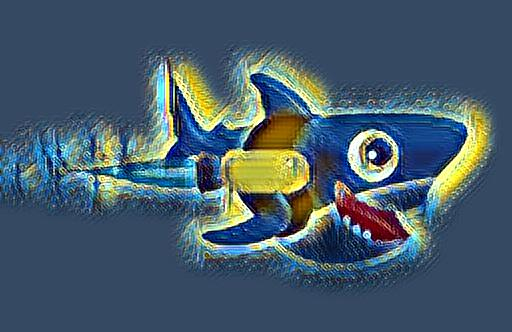## Prerequisites

To complete this tutorial, you will need:

Working with machine learning models can be memory intensive, so your machine should have at least 8GB of memory to perform some of the calculations in this tutorial.

## Step 1 — Installing Dependencies and Cloning the PyTorch-Style-Transfer GitHub Repository

In this tutorial, we’ll use an open-source implementation of neural style transfer provided by Hang Zhang called PyTorch-Style-Transfer. This particular implementation uses the `PyTorch` library.

Activate your programming environment, and install the `torchvision` and `torchfile` packages with the following command:

``````pip install torchvision torchfile

``````

Pip will automatically retrieve their dependencies:

``````OutputSuccessfully installed numpy-1.22.0 pillow-9.0.0 torch-1.10.1 torchfile-0.1.0 torchvision-0.11.2 typing-extensions-4.0.1
``````

To avoid cluttering your home directory with files, create a new directory called `style_transfer` and use it as your working directory:

``````mkdir style_transfer
cd style_transfer

``````

Next, clone the `PyTorch-Style-Transfer` repository to your working directory using the `git clone` command. You can learn more about Git in this Git tutorial series.

``````git clone https://github.com/zhanghang1989/PyTorch-Style-Transfer.git

``````

The author of this repository has placed the code we will be using in the `experiments` folder of the `PyTorch-Style-Transfer` repository, so switch to this directory once all files have been cloned:

``````cd PyTorch-Style-Transfer/experiments

``````

Take a look at the contents of the `experiments` directory:

``````ls

``````

You’ll see the following directories:

``````Outputcamera_demo.py  dataset  images  main.py  models  net.py  option.py  utils.py
``````

In this tutorial you’ll work with the `images/` directory, which contains stock images, and the `main.py` script, which is used to apply neural style transfer to your images.

Before moving to the next section, you also need to download the pre-trained deep learning model required to run neural style transfer. These models can be large and therefore not suitable for storing on GitHub, so the author provides a small script to download the file. You’ll find the script at `models/download_model.sh`.

First, make the script executable:

``````chmod +x ./models/download_model.sh

``````

``````./models/download_model.sh

``````

Now that everything’s downloaded, let’s use these tools to transform some images.

## Step 2 — Running your First Style Transfer Experiment

To illustrate how neural style transfer works, let’s start by using the example provided by the author of the `PyTorch-Style-Transfer` repository. Since we will need to display and view images, it will be more convenient to use a Jupyter notebook.

``````jupyter notebook

``````

Then access Jupyter by following the instructions presented.

Once Jupyter is displayed, create a new notebook by selecting New > Python 3 from the top right pull-down menu: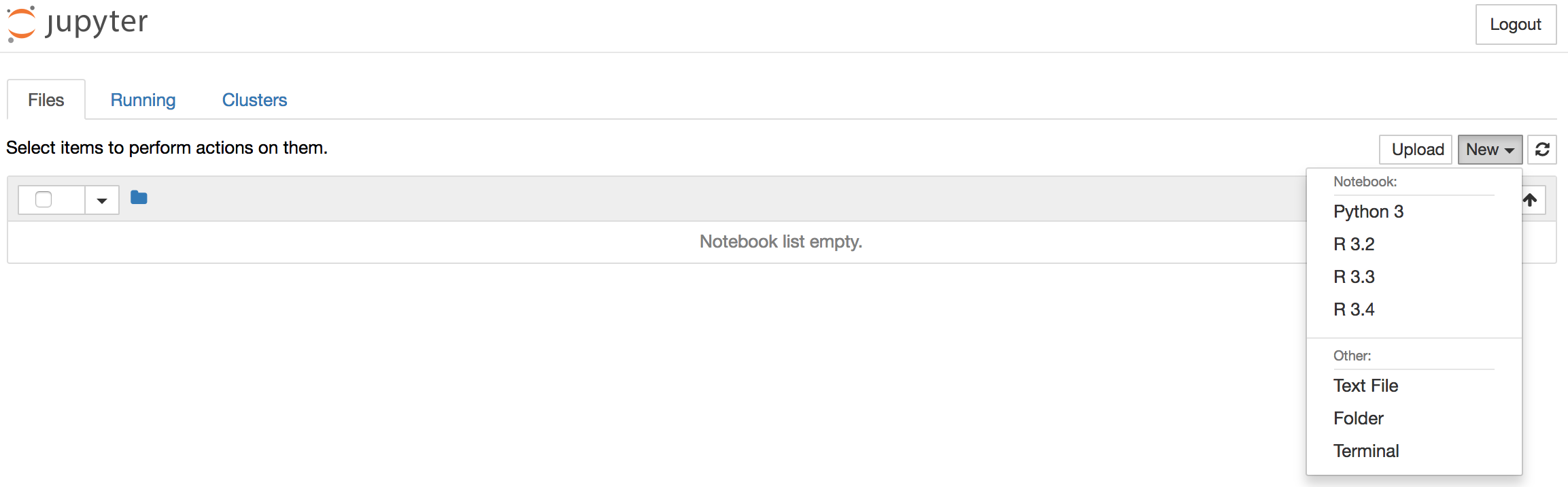This opens a new notebook where you can enter your code.

At the top of the notebook, add the following code to load the required libraries.

Notebook
``````import torch
import os
import subprocess
from IPython.display import Image
from IPython.display import display
``````

Along with `torch`, we’re also importing the standard libraries `os` and `subprocess`, which we’ll use to run Python scripts directly from Jupyter notebook. We also include the `IPython.display` library, which lets us display images within the Jupyter notebook.

Note: Type `ALT+ENTER` (or `SHIFT+ENTER` on macOS) to run the code and move into a new code block within your notebook. Do this after each code block in this tutorial to see your results.

The example provided in the `README` file of the `PyTorch-Style-Transfer` repository uses stock images located in the `images/` directory and the `main.py` script. You will need to provide at least five arguments in order to run the `main.py` script:

• The path to the content image (located in `/images/content`).
• The path to the style image (located in `/images/21styles`).
• The path to the pretrained GAN (Generative Adversarial Network) model used to perform the style transfer (located in `/models`).
• The path and name of the output image.
• Deep learning models run much faster on GPUs. If you have one available then specify the `--cuda=1` parameter, otherwise use `--cuda=0`.

To run the neural style transfer code, we’ll specify the required arguments and use the `subprocess` library to run the command in the shell.

First, let’s define the path to our working directory. We’ll store in a variable called `workingdir`:

Notebook
``````# define the path to the working directory
experiment_dir = 'style_transfer/PyTorch-Style-Transfer/experiments'
workingdir = '{}/{}'.format(os.environ['HOME'], experiment_dir)
``````

We’ll use this variable throughout our code when we point to images and other files.

Now let’s define the path to the `main.py` script, as well as the list of arguments that we will use as input for this test run. We’ll specify that the content image is `venice-boat.jpg`, the style image is`starry_night.jpg`, and we’ll save the output of our neural style transfer to a file called `test.jpg`:

Notebook
``````# specify the path to the main.py script
path2script = '{}/main.py'.format(workingdir)

# specify the list of arguments to be used as input to main.py
args = ['eval',
'--content-image',
'{}/images/content/venice-boat.jpg'.format(workingdir),
'--style-image',
'{}/images/21styles/starry_night.jpg'.format(workingdir),
'--model',
'{}/models/21styles.model'.format(workingdir),
'--output-image',
'{}/test.jpg'.format(workingdir),
'--cuda=0']
``````

Before running the test example, you can take a quick look at the content and style images that you have chosen for this example by executing this code in your notebook:

Notebook
``````content_image = Image('{}/images/content/venice-boat.jpg'.format(workingdir))
style_image = Image('{}/images/21styles/starry_night.jpg'.format(workingdir))
display(content_image)
display(style_image)
``````

You’ll see these images displayed in the output:Finally, concatenate the call to `main.py` and its list of arguments and run it in the shell using the `subprocess.check_output` function:

Notebook
``````# build subprocess command
cmd = ['python3', path2script] + args

# run the command
x = subprocess.check_output(cmd, universal_newlines=True)
``````

Depending on the amount of memory available on your machine, this may take a minute or two to run. Once it has completed, you should see a `test.jpg` file in your working directory. From a Jupyter notebook, you can use Ipython magic commands to display the contents of your working directory within the Jupyter notebook:

Notebook
``````!ls \$workingdir
``````

Alternatively, you can use the `ls` command in your terminal. Either way you’ll see the following output:

``````Output__pycache__	dataset  main.py  myutils  option.py
camera_demo.py	images	 models   net	   test.jpg
``````

You’ll see a new file called `test.jpg`, which contains the results of the neural style transfer using your input content and style images.

Use the `Image` function to display the content of `test.jpg`:

Notebook
``````Image('{}/test.jpg'.format(workingdir))
``````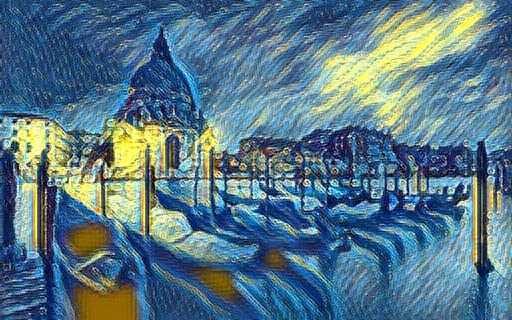The artistic style of Vincent van Vogh’s Starry Night canvas has been mapped to the content of our Venitian boat images. You’ve successfully applied neural style transfer with a textbook example, so let’s try repeating this exercise with different images.

## Step 3 — Transforming Your Own Images

So far, you’ve used the images provided by the author of the library we’re using. Let’s use our own images instead. To do this, you can either find an image you are interested in and use the URL for the image in the following command, or use the URL provided to use Sammy the Shark.

We’ll use some IPython magic again to download the image to our working directory and place it into a file called `sammy.png`.

Notebook
``````!wget -O - 'https://assets.digitalocean.com/blog/static/sammy-the-shark-gets-a-birthday-makeover-from-simon-oxley/sammy-jetpack.png' > \$workingdir/sammy.png
``````

When you run this command in your notebook, you’ll see the following output:

``````Output--2017-08-15 20:03:27--  https://assets.digitalocean.com/blog/static/sammy-the-shark-gets-a-birthday-makeover-from-simon-oxley/sammy-jetpack.png
Resolving assets.digitalocean.com (assets.digitalocean.com)... 151.101.20.233
Connecting to assets.digitalocean.com (assets.digitalocean.com)|151.101.20.233|:443... connected.
HTTP request sent, awaiting response... 200 OK
Length: 10483 (10K) [image/png]
Saving to: 'STDOUT'

-                   100%[===================>]  10.24K  --.-KB/s    in 0.001s

2017-08-15 20:03:27 (12.9 MB/s) - written to stdout [10483/10483]
``````

Use the `Image` command to display the new image in the notebook:

Notebook
``````Image('{}/sammy.png'.format(workingdir))
``````Following the same workflow as the test run, let’s run our artistic style transfer model using Rocket Sammy as the content image, and the same Starry Night picture as our style image.

We’ll use the same code we used previously, but this time we’ll specify the content image to be `sammy.png`, the style image to be `starry_night.jpg`, and we write the output to a file called `starry_sammy.jpg`. Then we execute the command:

Notebook
``````# specify the path to the main.py script
path2script = '{}/main.py'.format(workingdir)

# specify the list of arguments to be used as input to main.py
args = ['eval',
'--content-image',
'{}/sammy.png'.format(workingdir),
'--style-image',
'{}/images/21styles/starry_night.jpg'.format(workingdir),
'--model',
'{}/models/21styles.model'.format(workingdir),
'--output-image',
'{}/starry_sammy.jpg'.format(workingdir),
'--cuda=0']

# build subprocess command
cmd = ['python3', path2script] + args

# run the bash command
x = subprocess.check_output(cmd, universal_newlines=True)
``````

Then use the `Image` function to view the results of transferring the artistic style of Vincent van Vogh’s Starry Night to the content of your Rocket Sammy image.

Notebook
``````Image('{}/starry_sammy.jpg'.format(workingdir))
``````

You’ll see the new stylized Rocket Sammy:Let’s try this again by mapping a different style image to our picture of Rocket Sammy. We’ll use Picasso’s The Muse this time. Again, we use `sammy.png` as our content image, but we’ll change the style image to be `la_muse.jpg`. We’ll save the output to `musing_sammy.jpg`:

Notebook
``````# specify the path to the main.py script
path2script = '{}/main.py'.format(workingdir)

# specify the list of arguments to be used as input to main.py
args = ['eval',
'--content-image',
'{}/sammy.png'.format(workingdir),
'--style-image',
'{}/images/21styles/la_muse.jpg'.format(workingdir),
'--model',
'{}/models/21styles.model'.format(workingdir),
'--output-image',
'{}/musing_sammy.jpg'.format(workingdir),
'--cuda=0']

# build subprocess command
cmd = ['python3', path2script] + args

# run the bash command
x = subprocess.check_output(cmd, universal_newlines=True)
``````

Once the code has finished running, display the output of your work using the output filename you specified and the `Image` function:

Notebook
``````Image('{}/musing_sammy.jpg'.format(workingdir))
``````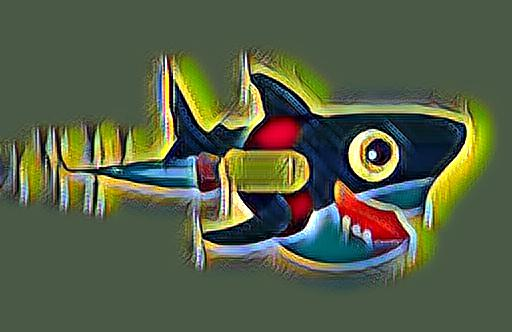By now, you should have a good idea how to use these transformations. Try using some of your own images if you haven’t already.

## Conclusion

In this tutorial, you used Python and an open-source PyTorch implementation of a neural style transfer model to apply stylistic transfer to images. The field of machine learning and AI is vast, and this is only one of its applications. Here are some additional things you can explore:

]]>
Thomas Vincent 2022-01-13T22:40:39.315Z
<![CDATA[Mobile app development company]]> tag:www.digitalocean.com,2005:/community/questions/mobile-app-development-company 2022-01-04T05:39:11.737Z Portraying the Silver Lining of Enterprise and Consumer-End Mobile Apps

]]>
startelelogic126 2022-01-04T05:39:11.737Z
<![CDATA[Coding for Beginners: Tutorials to Help New Developers]]> tag:www.digitalocean.com,2005:/community/tutorials/coding-for-beginners 2021-09-01T18:11:21.375Z Do you want to learn more technical skills but don’t know where to start? Explore our range of beginner-friendly tutorials to build your skills and launch your next project.

In this list, you’ll find tutorials on Developer Fundamentals, Programming Languages, Machine Learning, and Website Development.

## Developer Fundamentals

A Linux Command Line Primer The command-line interface is a powerful method for issuing commands to your computer or a remote cloud server. This tutorial introduces the command line with an interactive terminal you can launch in your browser.

How To Use SQL Structured Query Language — commonly known as SQL — is used to define, control, manipulate, and query data held in a relational database. SQL was first developed in the 1970s and is the predominant language used to manage relational database management systems today. Ideal for managing structured data (data that can fit neatly into an existing data model), SQL is an essential tool for developers and system administrators in a wide variety of contexts. Also, because of its maturity and prevalence, candidates with SQL experience are highly sought after for jobs across industries.

How To Deploy a Static Website to the Cloud with DigitalOcean App Platform If you’ve built a static website in a local environment, the next step is to decide how to publish it to the web. One way to publish your site is to deploy it as an application through App Platform, a DigitalOcean service that offers free hosting for three static sites. This tutorial will guide you through all the steps of deploying a static website to the cloud using App Platform, GitHub (a software development platform), and GitHub’s Desktop Application.

## Programming Languages

How To Code in JavaScript JavaScript, a high-level, object-based, dynamic scripting language, is a popular tool for making web pages interactive. Follow this tutorial series to learn JavaScript basics and create a “Hello, World” program.

How to Code in Python Python is a readable and versatile programming language used with applications ranging from web development to machine learning. It is an excellent tool for both new learners and experienced developers alike.

How To Code in React.js React is a popular JavaScript framework for creating front-end applications, such as user interfaces that allow users to interact with programs. Created by Facebook, it has gained popularity by enabling developers to create fast applications using an intuitive programming paradigm that ties JavaScript with an HTML-like syntax known as JSX.

How to Code in PHP PHP is a popular server scripting language known for creating dynamic and interactive web pages. Follow this tutorial series to learn the foundations of PHP, including how to create a “Hello, World” program.

How to Code in TypeScript TypeScript is an extension of the JavaScript language that uses JavaScript’s runtime with a compile-time type checker. This combination allows developers to use the full JavaScript ecosystem and language features while also providing optional static type-checking, enum data types, classes, and interfaces. This series will show you the syntax you need to get started with TypeScript, allowing you to leverage its typing system to make scalable, enterprise-grade code.

How to Code in Go Go (or GoLang) is a modern programming language originally developed by Google that uses high-level syntax similar to scripting languages. It is popular for its minimal syntax and innovative handling of concurrency, as well as for the tools it provides for building native binaries on foreign platforms.

## Machine Learning

How to Create an Intelligent Chatbot In this tutorial, you will create a chatbot that uses the OpenWeather API to tell the user the current weather in any city, and which is intelligent enough to communicate with the user in natural language. You will also learn the fundamentals of integrating a chatbot with an API.

An Introduction to Computer Vision OpenCV, or Open Source Computer Vision Library, is a powerful library used for image processing and image recognition. The library has a massive community and has been used extensively in many fields, from face detection to interactive art. It was first built in C++, but bindings have been created for different languages, such as Python and Java. In this project, you will create a web app that will allow users to detect circles in an uploaded image.

Introduction to PyTorch: Build a Neural Network to Recognize Handwritten Digits In this tutorial, you will build, train, and evaluate deep neural networks in PyTorch, a framework developed by Facebook AI Research for deep learning. When compared to other deep learning frameworks, like Tensorflow, PyTorch is a beginner-friendly framework with debugging features that aid in the building process. It’s also highly customizable for advanced users, with researchers and practitioners using it at companies like Facebook and Tesla.

How To Build a Neural Network to Translate Sign Language into English Computer vision is a subfield of computer science that aims to extract a higher-order understanding from images and videos. This powers technologies such as fun video chat filters, your mobile device’s face authenticator, and self-driving cars. In this tutorial, you’ll use computer vision to build an American Sign Language translator for your webcam. As you work through the tutorial, you’ll use OpenCV, a computer vision library. You will also use PyTorch to build a deep neural network and onnx to export your neural network.

## Website Development

How To Build a Website with HTML If you are interested in building and designing websites, HyperText Markup Language (HTML) is a great place to start. This project-based tutorial series will introduce you to HTML and its methods by building a personal website using our demonstration site as a model. After building your website, you can deploy it to the cloud on DigitalOcean’s App Platform for free.

How To Build a Website with CSS Cascading Style Sheets (CSS) is a stylesheet language used to control the presentation of websites. Alongside HTML and JavaScript, CSS is one of the core technologies of the World Wide Web. If you understand the fundamentals of HTML and are looking to grow your front-end development skills, learning CSS is a great next step. In this tutorial series, you will learn CSS by building a personal website.

How to Set Up Your First Gatsby Website Gatsby is a React framework that allows you to create static and serverless apps. Gatsby websites are different from traditional websites because they are usually deployed on a content delivery network (CDN) and are content-agnostic. The advantage of deploying from a CDN is that there is less latency and websites are usually served to the client faster.

How to Develop Websites with Vue.js This series provides a starting point for building websites with the front-end JavaScript framework Vue.js. Created in 2014 by Evan You (formally of Google), Vue.js is often described as a combination of React and Angular, borrowing the prop-driven development of React and the templating power of Angular. By the end of this series, you will have the tools to develop websites that focus on traditional HTML and CSS, while still taking advantage of the robustness and scalability of a front-end framework.

How To Install WordPress on Ubuntu 20.04 with a LAMP Stack WordPress is a popular open-source technology for creating websites and blogs. Used by 63% of all websites that use a content management system (CMS), WordPress sites represent 36% of all websites that are currently online. There are many different approaches to getting access to WordPress, and some setup processes are more complex than others. This tutorial is intended for those who desire to install and administer a WordPress instance on an unmanaged cloud server via the command line.

]]>
Erin Glass 2021-09-01T18:11:21.375Z
<![CDATA[How To Deploy a Pre-Trained Question and Answer TensorFlow.js Model on App Platform]]> tag:www.digitalocean.com,2005:/community/tutorials/how-to-deploy-a-pre-trained-question-and-answer-tensorflow-js-model-on-app-platform 2021-08-26T15:31:27.410Z The author selected Code 2040 to receive a donation as part of the Write for DOnations program.

### Introduction

As the field of machine learning (ML) grows, so does the list of environments for using this technology. One of these environments is the web browser, and in recent years, there has been a surge in data-related frameworks targeting web-based machine learning models. An example of these frameworks is TensorFlow.js, TensorFlow’s JavaScript counterpart library for training, executing, and deploying machine learning models in the browser. In an attempt to make TensorFlow.js accessible to developers with limited or no ML experience, the library comes with several pre-trained models that work out of the box.

A pre-trained machine learning model is ready-to-use machine learning you don’t have to train. TensorFlow.js includes 14 that suit a variety of use cases. For example, there is an image classifying model for identifying common objects and a body segmentation model for identifying body parts. The principal convenience of these models is that, as the name states, you don’t have to train them. Instead, you load them in your application. Besides their ease of use and pre-trained nature, these are curated models—they are accurate and fast, sometimes trained by the algorithms’ authors and optimized for the web browser.

In this tutorial, you will create a web application that serves a Question and Answer (QnA) pre-trained model using TensorFlow.js. The model you will deploy is a Bidirectional Encoder Representations from Transformers (BERT) model that uses a passage and a question as the input, and tries to answer the question from the passage.

You will deploy the app in DigitalOcean’s App Platform, a managed solution for building, deploying, and scaling applications within a few clicks from sources such as GitHub. The app you will create consists of a static page with two input fields, one for the passage and one for the question. In the end, you will have a written and deployed—from GitHub—a Question and Answer application using one of TensorFlow.js’ pre-trained models and DigitalOcean’s App Platform.

## Prerequisites

To complete this tutorial, you will need:

## Step 1 — Creating the App’s Interface and Importing the Required Libraries

In this step, you will write the app’s HTML code, which will define its interface and import the libraries for the app. The first of these libraries is TensorFlow.js, and instead of installing the package locally, you will load it from a content delivery network or CDN. A CDN is a network of servers spanning multiple locations that store content they provide to the internet. This content includes JavaScript files, such as the TensorFlow.js library, and loading it from a CDN saves you from packing them in your application. Similarly, you will import the library containing the Question and Answer model. In the next step, you will write the app’s JavaScript, which uses the model to answer a given question.

In this tutorial, you will structure an app that will look like this: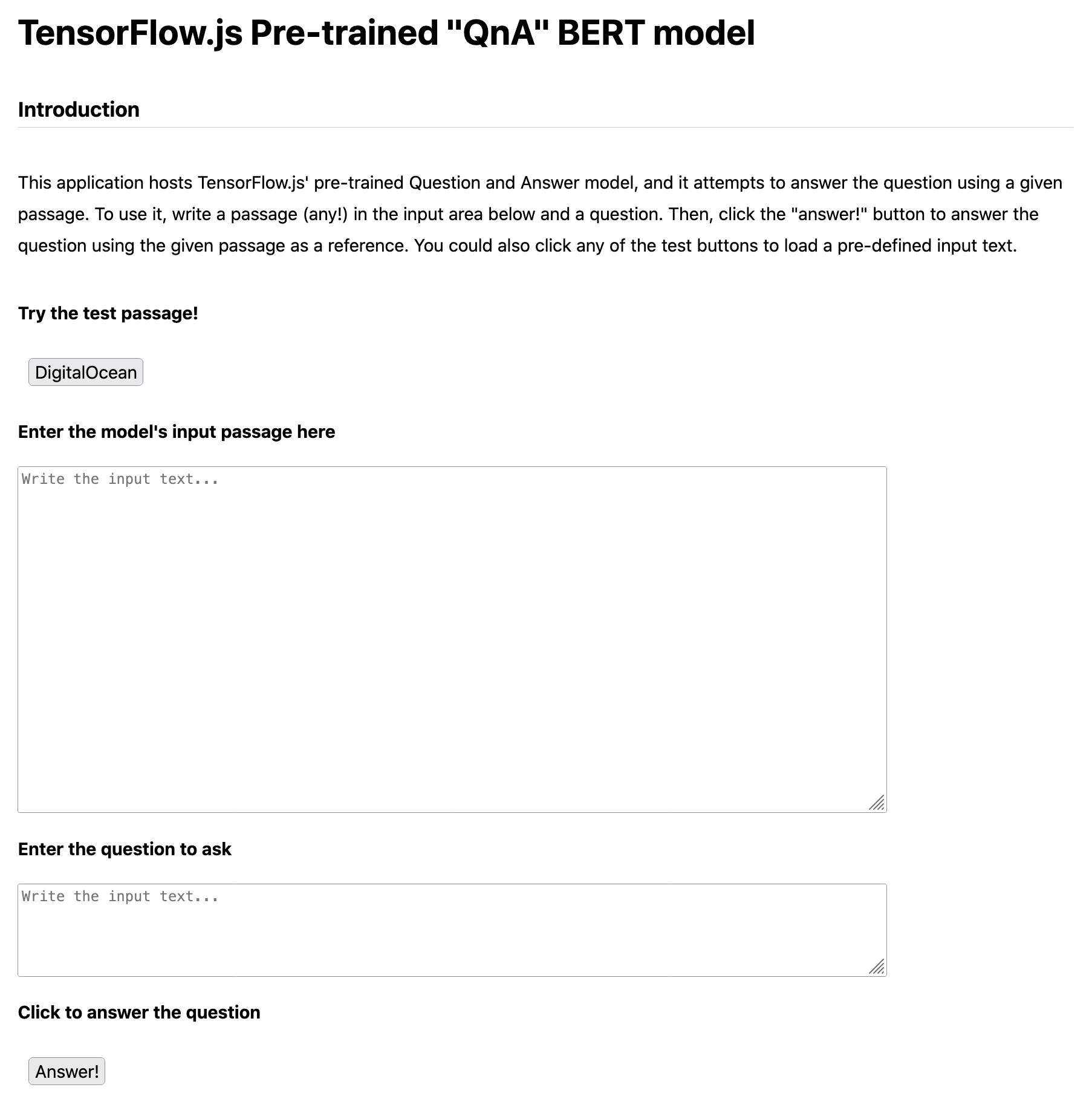The app has five major elements:

• A button that loads a pre-defined passage you can use to test the app.
• An input text field for a passage (if you choose to write or copy your own).
• An input text field for the question.
• A button that triggers the prediction that answers the question.
• An area to display the model’s output beneath the Answer! button (currently blank white space).

Start by creating a new directory named `tfjs-qna-do` at your preferred location. In this directory, using a text editor of your choice, create a new HTML file named `index.html` and paste in the following code:

index.html
``````<!DOCTYPE html>
<html lang="en-US">

<meta charset="utf-8" />
<script src="https://cdn.jsdelivr.net/npm/@tensorflow/tfjs"></script>
<!-- Load the QnA model -->
<script src="https://cdn.jsdelivr.net/npm/@tensorflow-models/qna"></script>

<body>
<div class="main-centered-container">
<h1>TensorFlow.js Pre-trained "QnA" BERT model</h1>
<p>This application hosts TensorFlow.js' pre-trained Question and Answer model, and it attempts to answer the question
using a given passage. To use it, write a passage (any!) in the input area below and a question. Then, click the
"answer!" button to answer the question using the given passage as a reference. You could also click the test
button to load a pre-defined input text.</p>

<h4>Try the test passage!</h4>
<div id='test-buttons'></div>

<div>
<h4>Enter the model's input passage here</h4>
<textarea id='input-text' rows="20" cols="100" placeholder="Write the input text..."></textarea>
</div>

<div>
<textarea id='question' rows="5" cols="100" placeholder="Write the input text..."></textarea>
</div>

<script src="./index.js"></script>

</div>
</body>

</html>
``````

Here’s how that HTML breaks down:

• The initial `<html>` tag has the `<head>` tag that’s used for defining metadata, styles, and loading scripts. Its first element is `<meta>`, and here you will set the page’s `charset` encoding to `utf-8`. After it, there are two `<script>` tags for loading both TensorFlow.js and the Question and Answer model from a CDN.
• Following the two `<script>` tags, there’s a `<link>` tag that loads a CSS file (which you will create next).
• Next, there’s the HTML’s `<body>`—the document’s content. Inside of it, there’s a `<div>` tag of class `main-centered-container` containing the page’s elements. The first is a `<h1>` header with the application title and a smaller `<h3>` header, followed by a brief introduction explaining how it works.
• Under the introduction, there’s a `<h4>` header and a `<div>` where you will append buttons that populate the passage input text field with sample text.
• Then, there are the app’s input fields: one for the passage (what you want the model to read) and one for the question (what you want the model to answer). If you wish to resize them, change the `rows` and `cols` attributes.
• After the text fields, there’s a `<div>` with id `button` where you will later append a button that, upon clicking, reads the text fields’ text and uses them as an input to the model.
• Last, there is a `<div>` with id `answer` that’s used to display the model’s output and a `<script>` tag to include the JavaScript code you will write in the following section.

Next, you’ll add CSS to the project. In the same directory where you added the `index.html` file, create a new file named `style.css` and add the following code:

style.css
``````body {
margin: 50px 0;
font-family: -apple-system, BlinkMacSystemFont, "Segoe UI", Roboto, Helvetica, Arial;
}

button {
margin: 10px 10px;
font-size: 100%;
}

p {
line-height: 1.8;
}

.main-centered-container {
display: flex;
flex-direction: column;
margin: 0 auto;
max-width: 960px;
}

border: solid #d0d0d0;
border-width: 0 0 1px;
}
``````

This CSS adds style to three HTML elements: body, buttons, and paragraphs (`<p>`). To `body`, it adds a margin, padding, and changes the default font. To `button`, it adds margin and increases the font size. To paragraph `p`, it modifies the `line-height` attribute. The CSS has a class `main-centered-container` that centers the content and another one, `header-border`, that adds a solid line to an element.

In this step, you wrote the app’s HTML file. You imported the TensorFlow.js library and QnA model, and defined the elements you will use later to add the passage and the question you want to answer. In the following step, you will write the JavaScript code that reads the elements and triggers the predictions.

## Step 2 — Predicting with the Pre-Trained Model

In this section, you will implement the web app’s JavaScript code that reads the app’s input fields, makes a prediction, and writes the predicted answers in HTML. You will do it in one function that’s triggered when you click the “answer” button. Once clicked, it will reference both input fields to get their values, use them as inputs to the model, and write its output in the `<div>` with id `output` you defined in Step 1. Then, you will run the app locally to test it before adding it to GitHub.

In the project’s directory `tfjs-qna-do/`, create a new file named `index.js`, and declare the following variables:

index.js
``````let model;

// The text field containing the input text
let inputText;

// The text field containing the question
let questionText;

// The div where we will write the model's answer
``````

The first of the variables, `model`, is where you will store the QnA model; `inputText` and `questionText` are references to the input and question text fields; `answersOutput` is a reference to the output `<div>`.

In addition to these variables, you will need a constant for storing the sample text you will use to test the app. We will use the Wikipedia article on DigitalOcean as a sample passage. Based on this passage, you could ask the model questions like “Where is DigitalOcean headquartered?” and hopefully, it will output “New York”.

Copy this block into your `index.js` file:

index.js
``````// Sample passage from Wikipedia.
const doText = `DigitalOcean, Inc. is an American cloud infrastructure provider headquartered in New York City with data centers worldwide.
DigitalOcean provides developers cloud services that help to deploy and scale applications that run simultaneously on multiple computers.
DigitalOcean also runs Hacktoberfest which is a month-long celebration (October 1-31) of open source software run in partnership with GitHub and Twilio.
`;
``````

Now, you will define the app’s functions, starting with `createButton()`. This function creates a button and appends it to an HTML element:

index.js
``````function createButton(innerText, id, listener, selector, disabled = false) {
const btn = document.createElement('BUTTON');
btn.innerText = innerText;
btn.id = id;
btn.disabled = disabled;

document.querySelector(selector).appendChild(btn);
}
``````

`createButton()` is a function that creates the app’s two buttons; since all the buttons work similarly, this function avoids repeating code. The function has five parameters:

• `innerText`: the button’s text.
• `id`: the button’s id.
• `listener`: a callback function that’s executed when the user clicks the button.
• `selector`: the `<div>` element where you will append the button.
• `disabled`: a boolean value to disable or enable the button. This parameter’s default value is `false`.

`createButton()` starts by creating an instance of a button and assigns it to the variable `btn`. Then, it sets the button’s `innerText`, `id`, and `disabled` attributes. The button uses a click event listener that executes a callback function whenever you click it. The function’s last line appends the button to the `<div>` element specified in the `selector` parameter.

Next, you will create a new function named `setupButtons()` that calls `createButton()` two times to create the app’s buttons. Begin by creating the app’s Answer! button:

index.js
``````function setupButtons() {
// Button to predict
() => {
// Write the answers to the output div as an unordered list.
// It uses map create a new list of the answers while adding the list tags.
// Then, we use join to concatenate the answers as an array with a line break
.join('<br>');

}).catch((e) => console.log(e));
}
``````

The first button the function creates is the one that triggers the predictions. Its first two arguments, `innerText` and `id`, are the button’s text and the identifier you want to assign to the button. The third argument is the listener’s callback (explained below). The fourth argument, `selector`, is the id of the <div> where you want to add the button (`#answer-button`), and the fifth argument, `disabled`, is set to `true`, which disables the button (to avoid predicting before the app loads the model).

The listener’s callback is a function that’s executed once you click the Answer! button. Once clicked, the callback function first calls the pre-trained model’s function `findAnswers()`. (For more about the `findAnswers()` function, see the product documentation). `findAnswers()` uses as arguments the input passage (read from `questionText`) and the question (read from `inputText`). It returns the model’s output in an array that looks as follows:

``````Model's output[
{
"text": "New York City",
"score": 19.08431625366211,
"startIndex": 84,
"endIndex": 97
},
{
"text": "in New York City",
"score": 8.737937569618225,
"startIndex": 81,
"endIndex": 97
},
{
"text": "New York",
"score": 7.998648166656494,
"startIndex": 84,
"endIndex": 92
},
{
"text": "York City",
"score": 7.5290607213974,
"startIndex": 88,
"endIndex": 97
},
{
"text": "headquartered in New York City",
"score": 6.888534069061279,
"startIndex": 67,
"endIndex": 97
}
]
``````

Each of the array’s elements is an object of four attributes:

• `text`: the answer.
• `score`: the model confidence level.
• `startIndex`: the index of the passage’s first character that answers the question.
• `endIndex`: the index of the answer’s last characters.

Instead of displaying the output as the model returns it, the callback uses a `map()` function that creates a new array containing the model’s answers and scores while adding the list `<li>` HTML tags. Then, it joins the array’s elements with a `<br>` (line break) between answers and assigns the result to the `answer` `<div>` to display them as a list.

Next, add a second call to `createButton()`. Add the highlighted portion to the `setupButtons` function beneath the first `createButton`:

index.js
``````function setupButtons() {
// Button to predict
() => {
// Write the answers to the output div as an unordered list.
// It uses map create a new list of the answers while adding the list tags.
// Then, we use join to concatenate the answers as an array with a line break
.join('<br>');

}).catch((e) => console.log(e));

createButton('DigitalOcean', 'test-case-do-btn',
() => {
document.getElementById('input-text').value = doText;
}, '#test-buttons', false);
}
``````

This new call appends to the `test-buttons` `<div>` the button that loads the DigitalOcean sample text you defined earlier in the variable `doText`. The function’s first argument is the label for the button (DigitalOcean), the second argument is the `id`, the third is the listener’s callback that writes in the passage input text area the value of the `doText` variable, the fourth is the `selector`, and the last is a `false` value (to avoid disabling the button).

Next, you will create a function, named `init()`, that calls the other functions:

index.js
``````async function init() {
setupButtons();
inputText = document.getElementById('input-text');
questionText = document.getElementById('question');

}
``````

`init()` starts by calling `setupButtons()`. Then, it assigns some of the app’s HTML elements to the variables you defined at the top of the script. Next, it loads the QnA model and changes to `false` the disabled attribute of the answer (`answer-btn`) button.

Last, call `init()`:

index.js
``````init();
``````

You have finished the app. To test it, open your web browser and write the project’s directory absolute path with `/index.html` appended to it in the address bar. You could also open your file manager application (such as Finder on Mac) and click on “index.html” to open the web application. In this case, the address would look like `your_filepath/tfjs-qna-do/index.html`.

To find the absolute path, go to the terminal and execute the following command from the project’s directory:

``````pwd

``````

Its output will look like this:

``````Output/Users/your_filepath/tfjs-qna-do
``````

After launching the app, you will need to wait a few seconds while it downloads and loads the model. You will know it’s ready when the app enables the Answer! button. Then, you could either use the test passage button (`DigitalOcean`) or write your own passage, and then input a question.

To test the app, click the “DigitalOcean” button. In the passage input area, you will see the sample text about DigitalOcean. In the question input area, write “Where is DigitalOcean headquartered?” From the passage, we can see the answer is “New York”. But will the model say the same? Click Answer! to find out.

The output should look similar to this: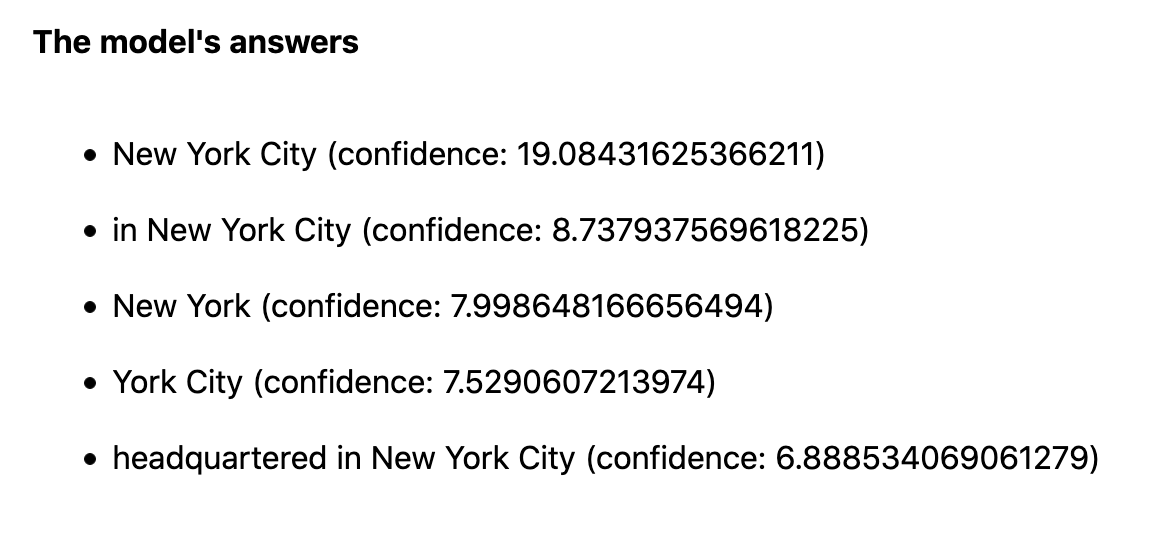Correct! Although there are slight differences, four of the five answers say that DigitalOcean’s headquarters are in New York City.

In this step, you completed and tested the web application. The JavaScript code you wrote reads the input passage and question and uses it as an input to the QnA model to answer the question. Next, you will add the code to GitHub.

## Step 3 — Pushing the App to GitHub

In this section, you will add the web application to a GitHub repository. Later, you will connect the repository to DigitalOcean’s App Platform and deploy the app.

Start by logging in to GitHub. From its main page, click the green button under your name or the plus sign on the screen’s upper right corner to create a new repository.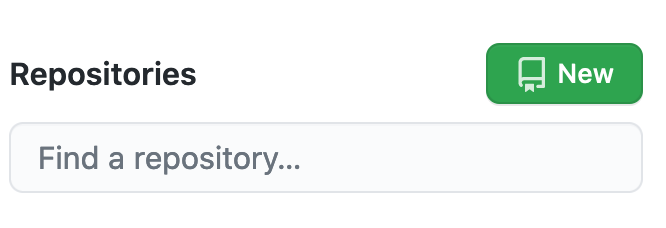Either choice takes you to the Create a new repository screen. In the Repository name field (after your username), name the repository tfjs-qna-do. Select its privacy setting, and click Create repository to create it.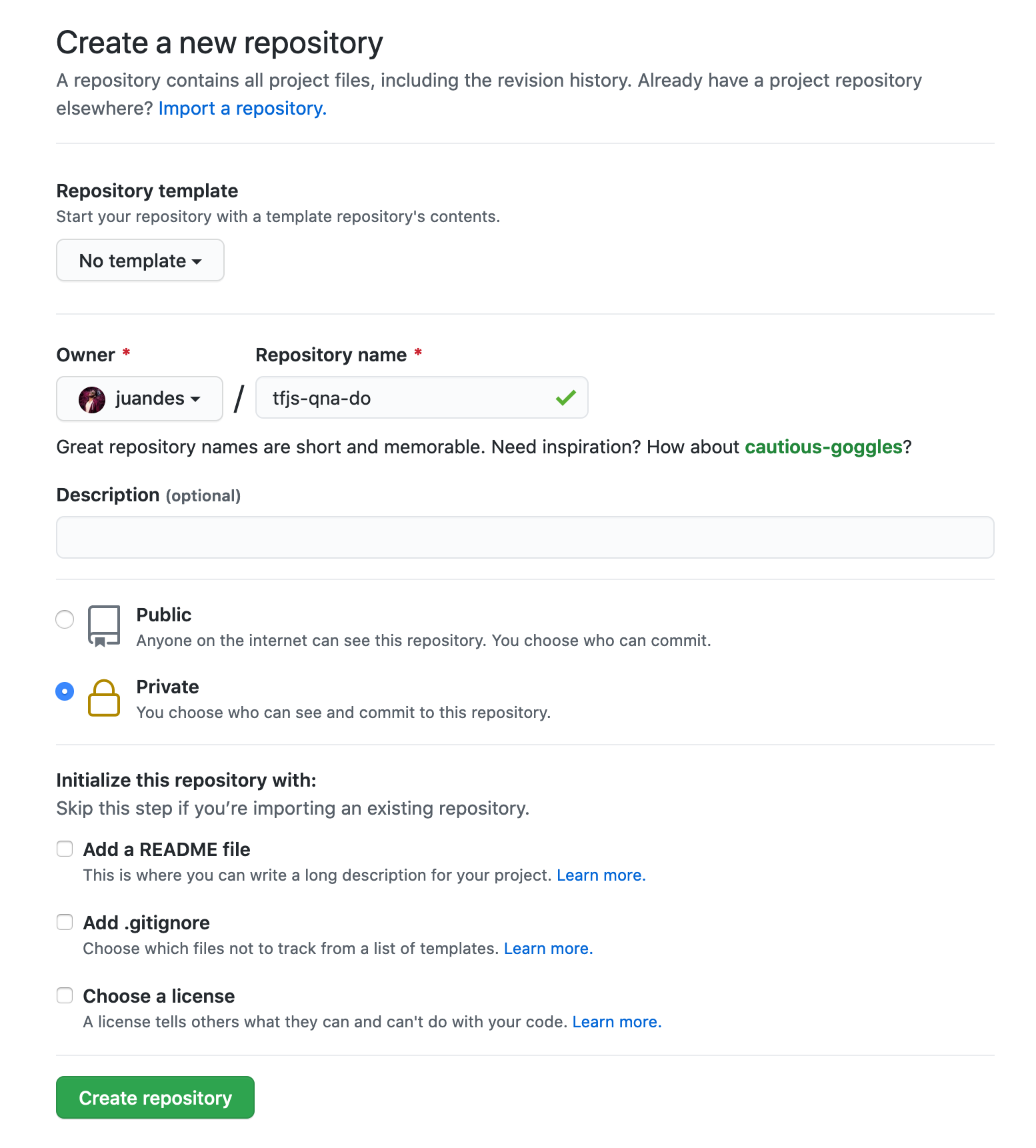Open a terminal and go to the project’s directory `tfjs-qna-do/`. There, execute the following command to create a new local Git repository:

``````git init

``````

Next, stage the files you want to track with Git, which in this case, is everything in the directory:

``````git add .

``````

And commit them:

``````git commit -m "initial version of the app"

``````

Rename the repository’s principal branch to `main`:

``````git branch -M main

``````

``````git remote add origin git@github.com:your-github-username/tfjs-qna-do.git

``````

Last, push the local codebase to the remote repository:

``````git push -u origin main

``````

It will ask you to enter your GitHub credentials if this is the first time you are pushing code to it.

Once you have pushed the code, return to the GitHub repository and refresh the page. You should see your code.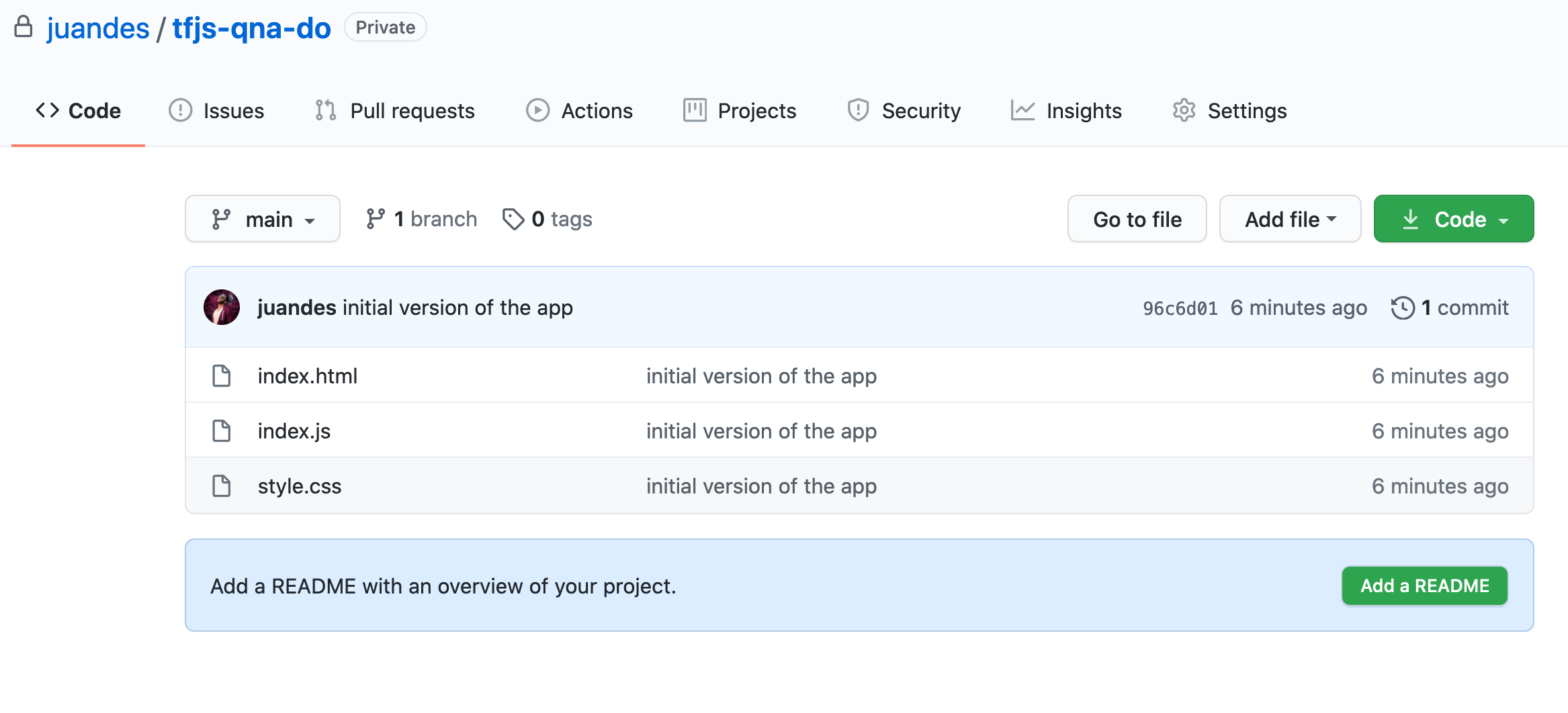In this step, you have pushed your web app’s code to a remote GitHub repository. Next, you will link this repository to your DigitalOcean account and deploy the app.

## Step 4 — Deploying the Web Application in DigitalOcean App Platform

In this last section, you will deploy the Question and Answer app to DigitalOcean’s App Platform) from the GitHub repository created in Step 3.

Start by logging into your DigitalOcean account. Once logged in, click the Create green button in the upper right corner and then on Apps; this takes you to the Create new app screen: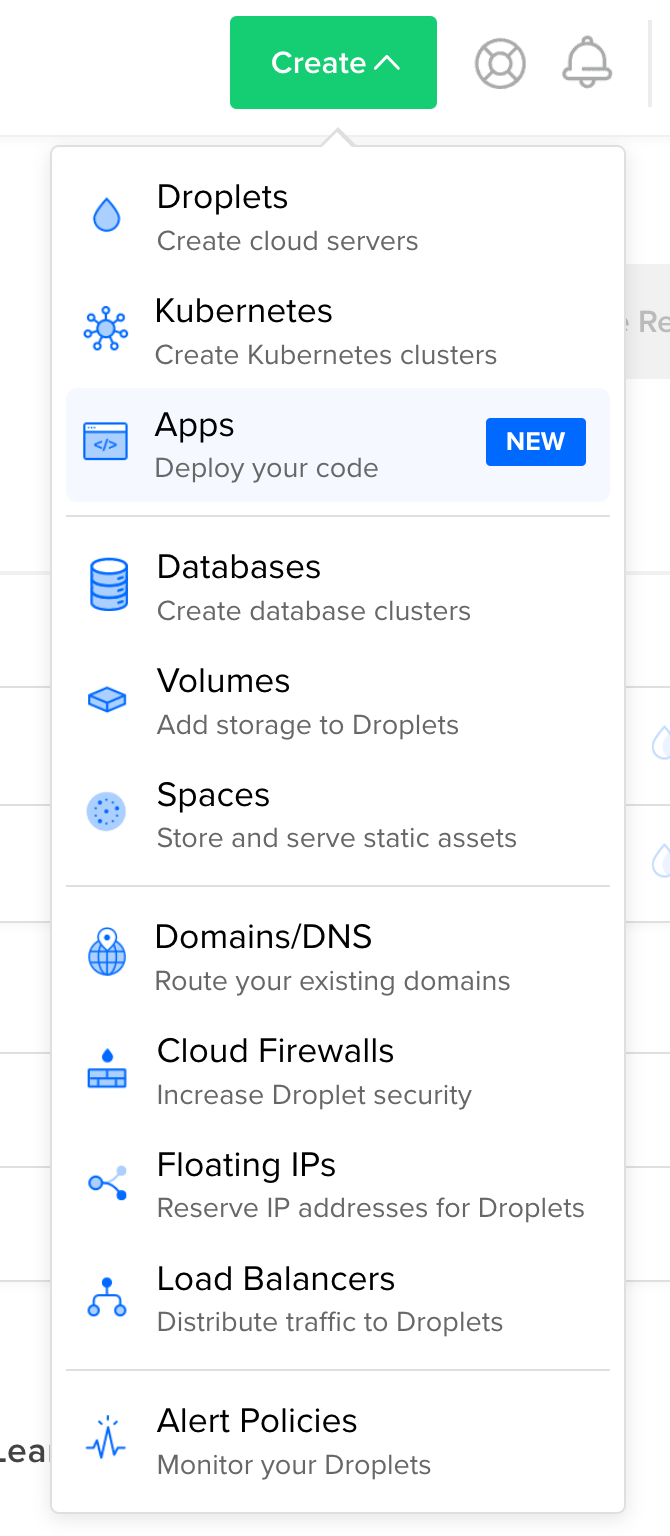Here, choose GitHub as the source where the project resides.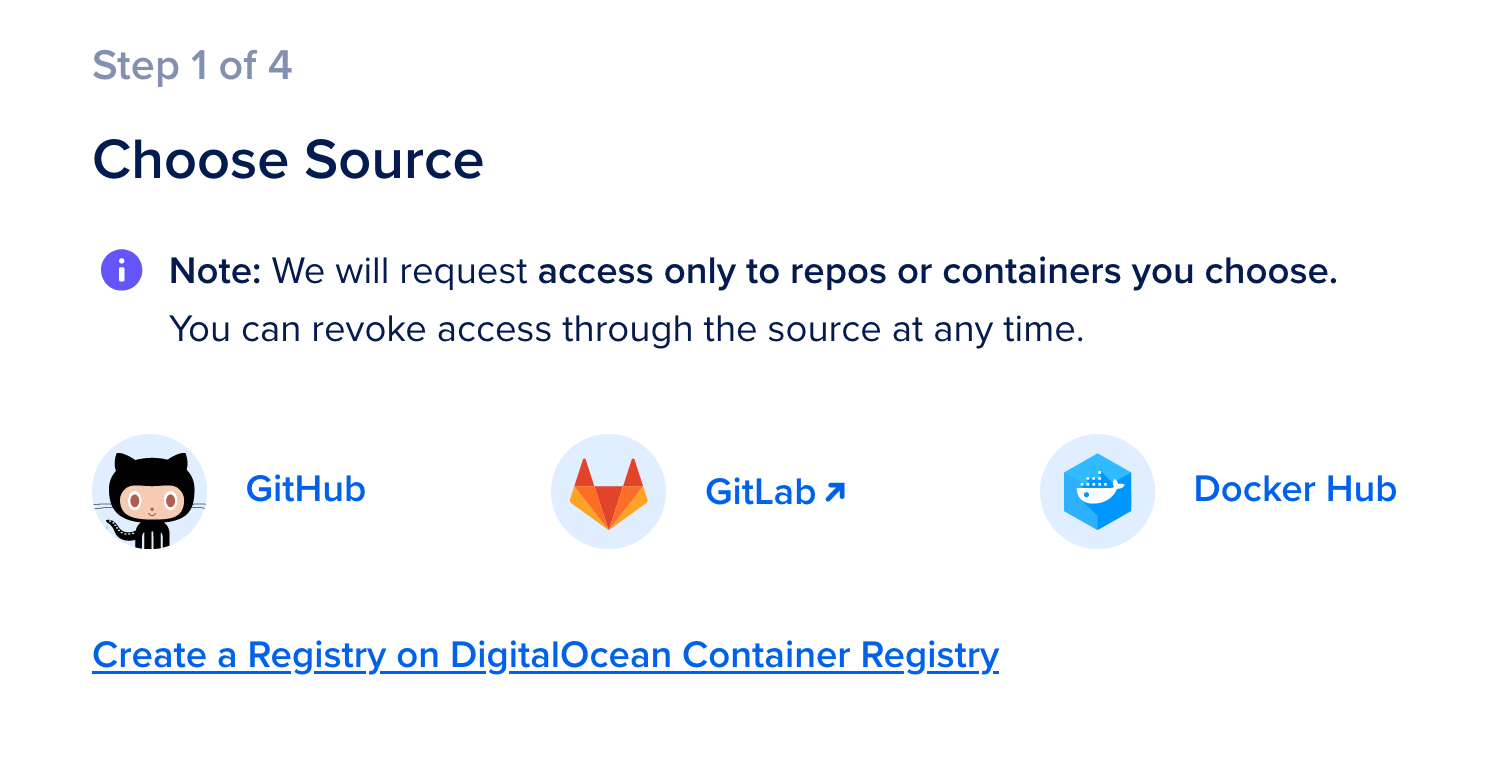If you haven’t linked your DigitalOcean account with GitHub, it will ask you to authorize DigitalOcean to access your GitHub. After doing so, select the repository you want to link: tfjs-qna-do.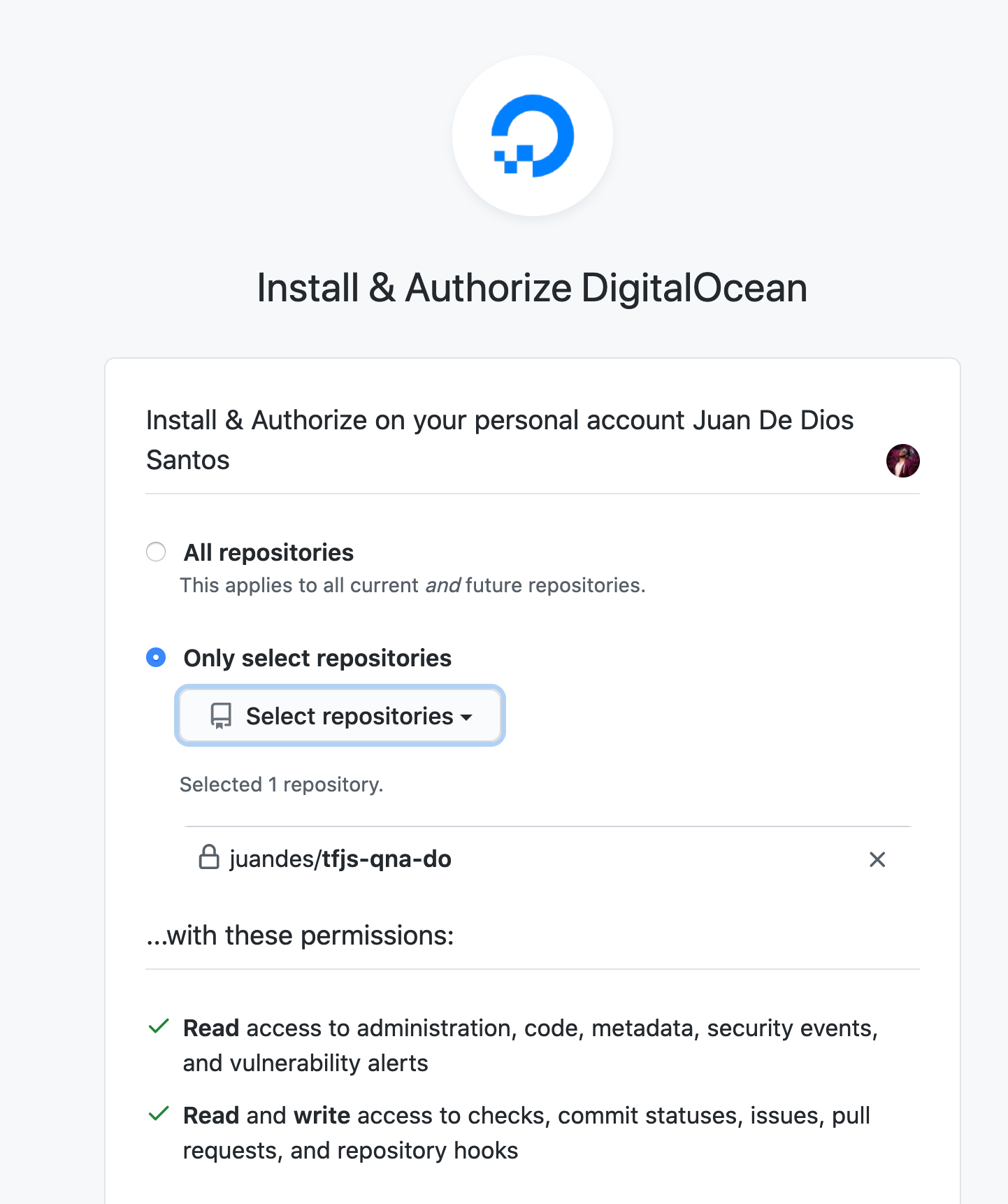Back on the App Platform window, select the app’s repository (tfjs-qna-do), the branch from where it will deploy the app (main), and check the Autodeploy code changes box; this option ensures that the application gets re-deployed every time you push code to the main branch.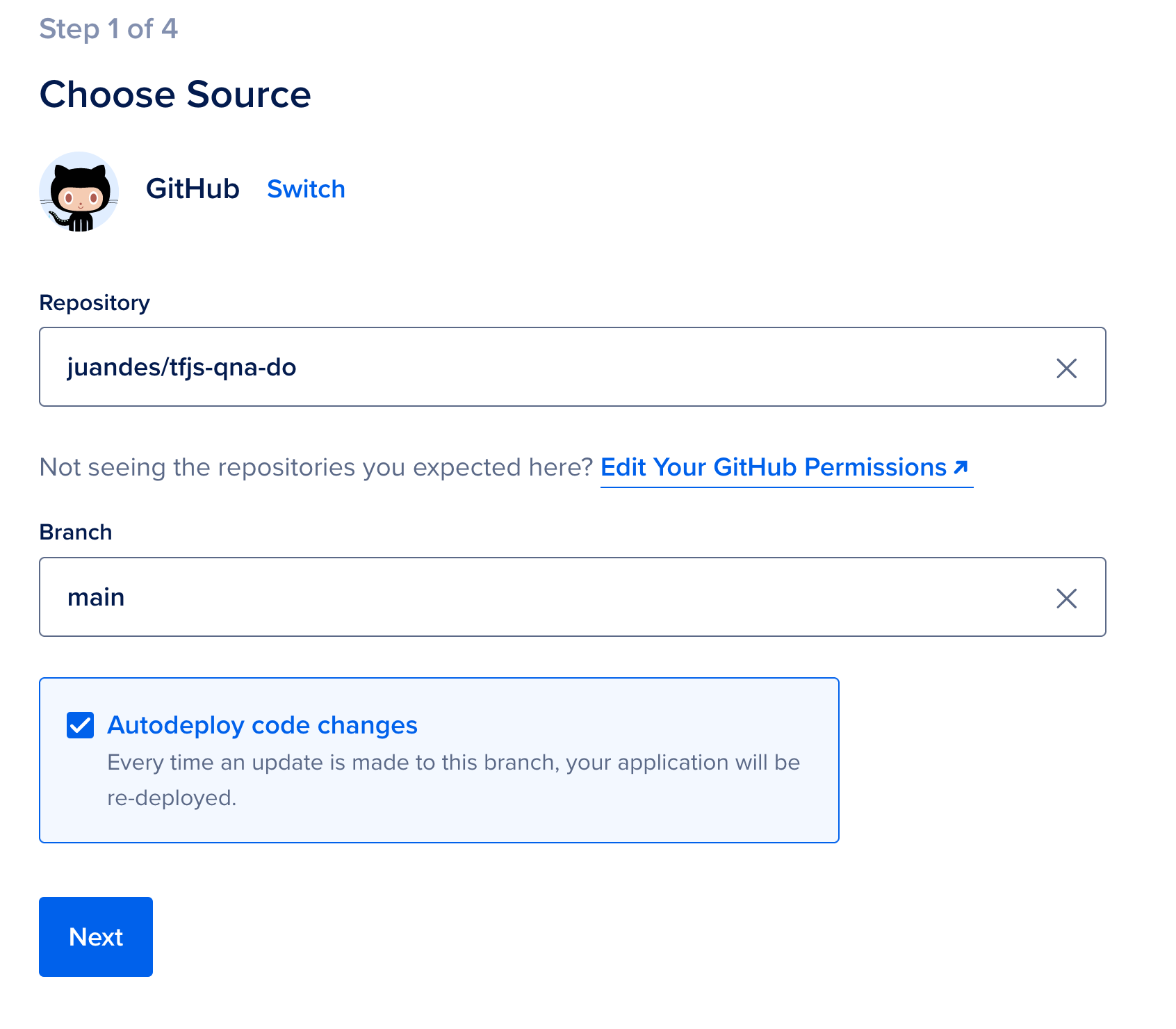In the next screen, Configure your app, use the default configuration values. As an additional step, if you wish to change the web app’s HTTP requests route, from, for example, the default https://example.ondigitalocean.app/tfjs-qna-do, to https://example.ondigitalocean.app/my-custom-route, click on Edit under HTTP Routes and write my-custom-route in the ROUTES input.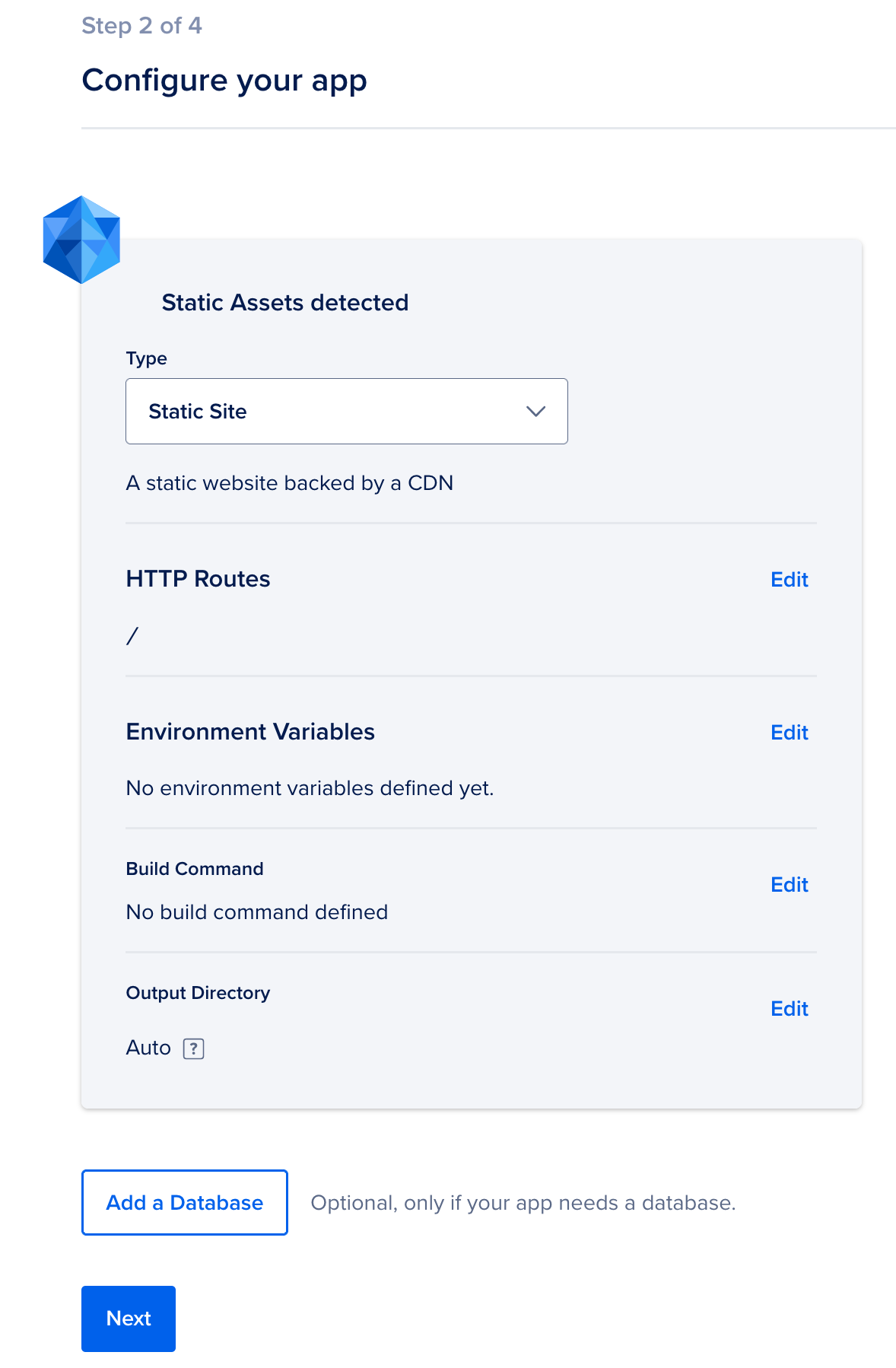Next, you will name the site. Again, you could leave the default tfjs-qna-do name or replace it with another one.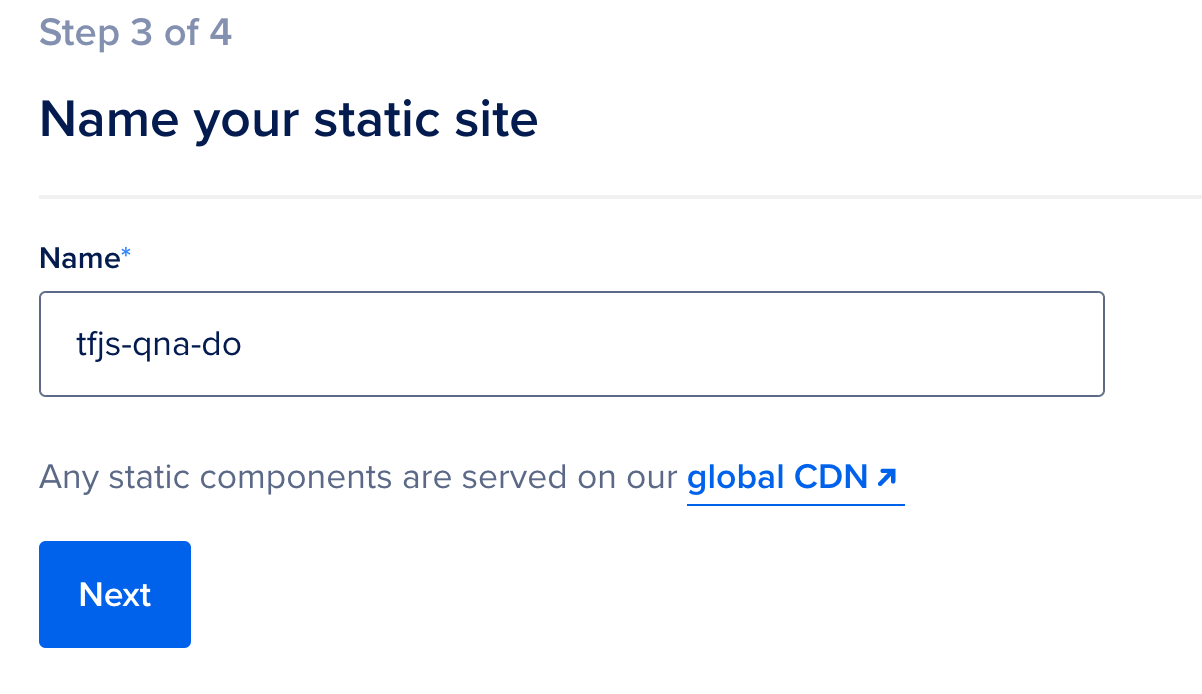Clicking Next will take you to the last screen, Finalize and launch, where you will select the app’s pricing tier. Since the app is a static webpage, App Platform will automatically select the free Starter plan. Last, click the Launch Starter App button to build and deploy the application.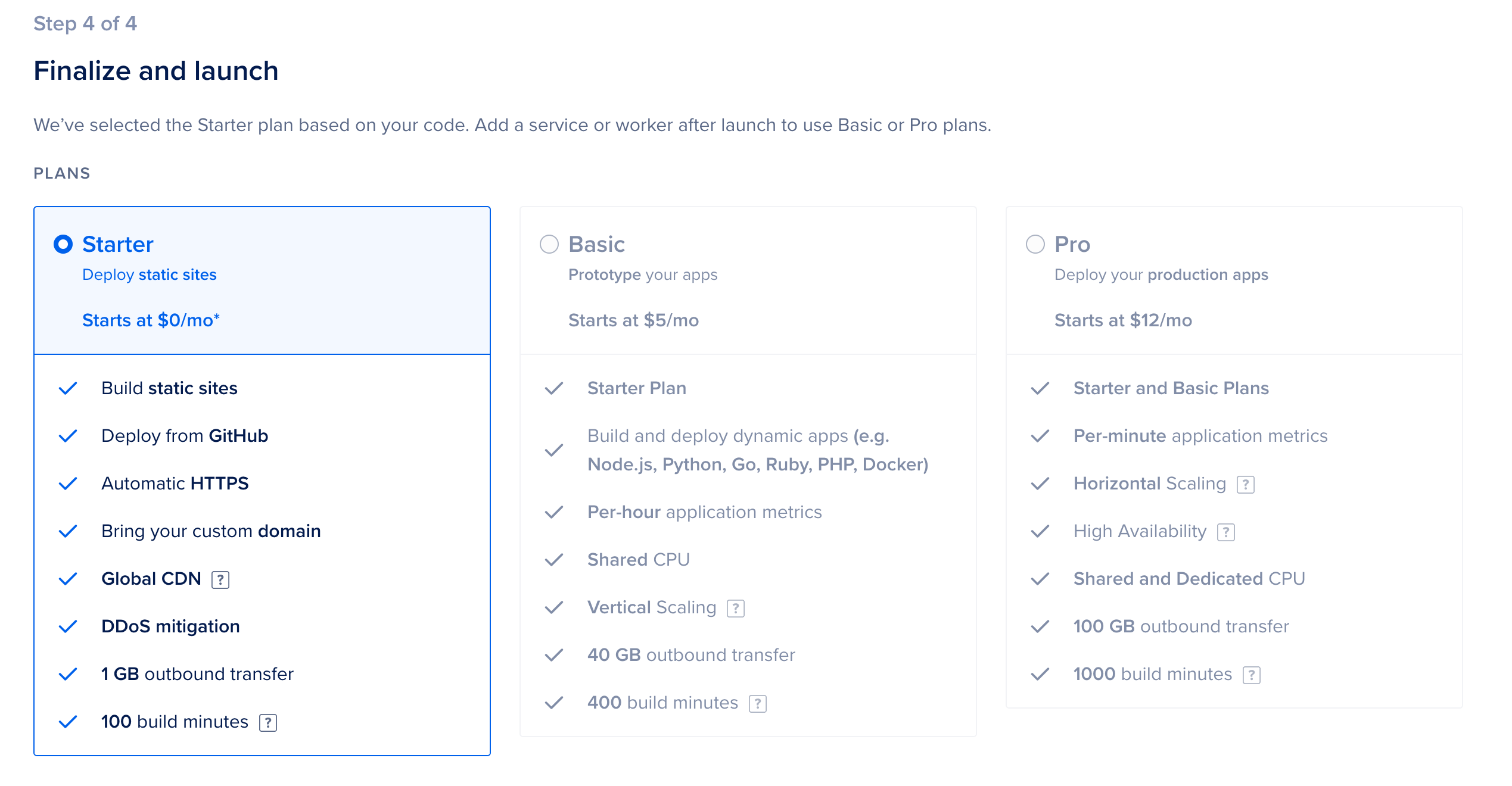While the app is deployed, you will see a screen similar to this: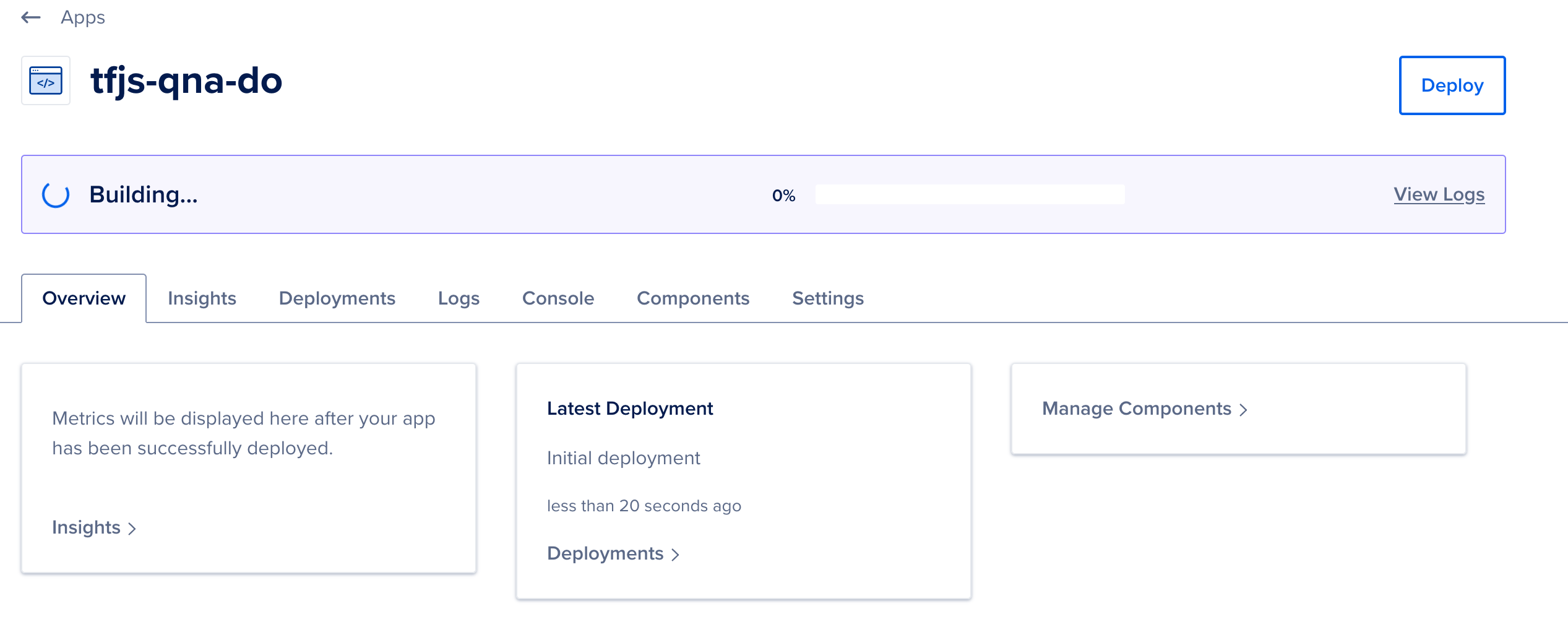And once deployed, you will see: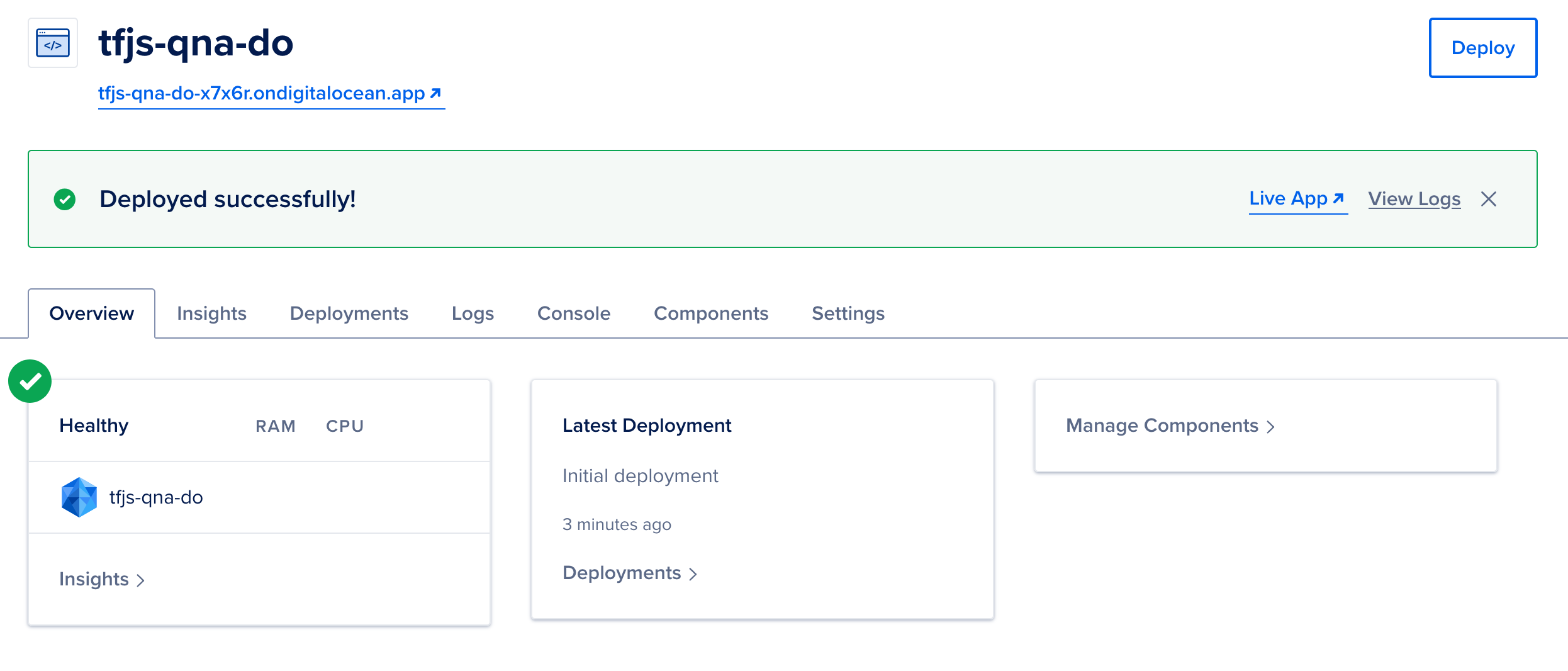To access the app, click on the app’s URL to access your app, which is now deployed in the cloud.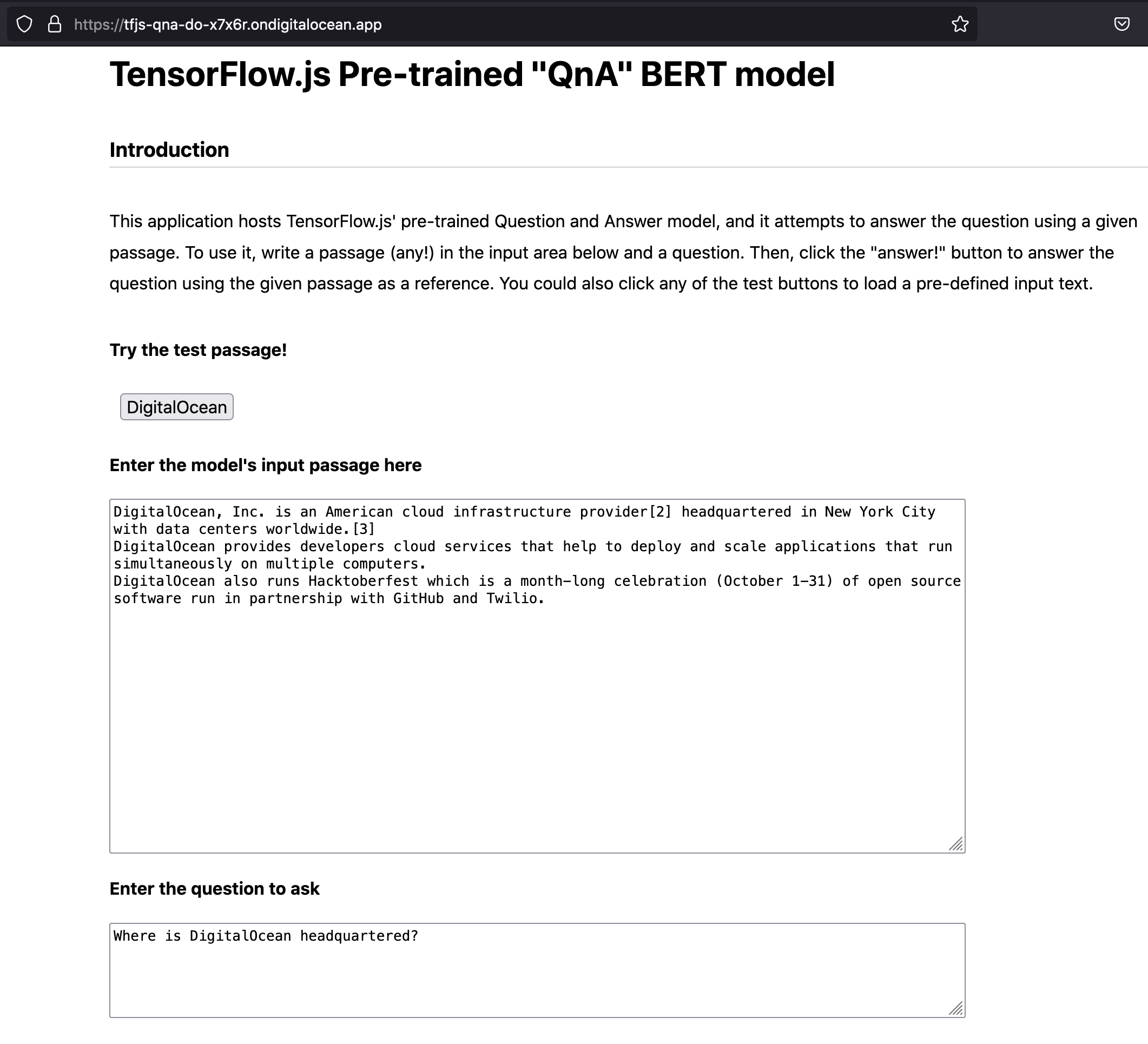## Conclusion

As the field of machine learning expands, so do its use cases alongside the environments and platforms it reaches – including the web browser.

In this tutorial, you have built and deployed a web application that uses a TensorFlow.js pre-trained model. Your Question and Answer web app takes as input a passage along with a question and uses a pre-trained BERT model to answer the question according to the passage. After developing the app, you linked your GitHub account with DigitalOcean and deployed the application in App Platform without needing additional code.

As future work, you could trigger the app’s automatic deployment by adding a change to it and pushing it to GitHub. You could also add a new test passage and format the answer’s output as a table to improve its readability.

]]>
Juan De Dios Santos Rivera 2021-08-26T15:31:27.410Z
<![CDATA[How To Build a Neural Network to Recognize Handwritten Digits with TensorFlow]]> tag:www.digitalocean.com,2005:/community/tutorials/how-to-build-a-neural-network-to-recognize-handwritten-digits-with-tensorflow 2021-08-11T20:37:47.451Z Introduction

Neural networks are used as a method of deep learning, one of the many subfields of artificial intelligence. They were first proposed around 70 years ago as an attempt at simulating the way the human brain works, though in a much more simplified form. Individual ‘neurons’ are connected in layers, with weights assigned to determine how the neuron responds when signals are propagated through the network. Previously, neural networks were limited in the number of neurons they were able to simulate, and therefore the complexity of learning they could achieve. But in recent years, due to advancements in hardware development, we have been able to build very deep networks, and train them on enormous datasets to achieve breakthroughs in machine intelligence.

These breakthroughs have allowed machines to match and exceed the capabilities of humans at performing certain tasks. One such task is object recognition. Though machines have historically been unable to match human vision, recent advances in deep learning have made it possible to build neural networks which can recognize objects, faces, text, and even emotions.

In this tutorial, you will implement a small subsection of object recognition—digit recognition. Using TensorFlow, an open-source Python library developed by the Google Brain labs for deep learning research, you will take hand-drawn images of the numbers 0-9 and build and train a neural network to recognize and predict the correct label for the digit displayed.

While you won’t need prior experience in practical deep learning or TensorFlow to follow along with this tutorial, we’ll assume some familiarity with machine learning terms and concepts such as training and testing, features and labels, optimization, and evaluation. You can learn more about these concepts in An Introduction to Machine Learning.

## Prerequisites

To complete this tutorial, you’ll need:

Note: TensorFlow requires a minimum Python version of 3.5 and supports versions up to 3.8. Older or newer versions of Python are not supported. This tutorial is designed to work with TensorFlow versions 1.4.0-1.15.5 with Python 3.6. Newer or older version of either will likely result in installation or runtime errors. For a full list of version requirements for Windows, macOS, and Linux, visit the Install TensorFlow with pip page on the TensorFlow site.

## Step 1 — Configuring the Project

Before you can develop the recognition program, you’ll need to install a few dependencies and create a workspace to hold your files.

We’ll use a Python 3 virtual environment to manage our project’s dependencies. Create a new directory for your project and navigate to the new directory:

``````mkdir tensorflow-demo
cd tensorflow-demo

``````

Execute the following commands to set up the virtual environment for this tutorial:

``````python3 -m venv tensorflow-demo
source tensorflow-demo/bin/activate

``````

Next, install the libraries you’ll use in this tutorial. We’ll use specific versions of these libraries by creating a `requirements.txt` file in the project directory which specifies the requirement and the version we need. Create the `requirements.txt` file:

``````touch requirements.txt

``````

Open the file in your text editor and add the following lines to specify the Image, NumPy, and TensorFlow libraries and their versions:

``````requirements.txtimage==1.5.20
numpy==1.14.3
tensorflow==1.4.0
``````

Save the file and exit the editor. Then install these libraries with the following command:

``````pip install -r requirements.txt

``````

With the dependencies installed, we can start working on our project.

## Step 2 — Importing the MNIST Dataset

The dataset we will be using in this tutorial is called the MNIST dataset, and it is a classic in the machine learning community. This dataset is made up of images of handwritten digits, 28x28 pixels in size. Here are some examples of the digits included in the dataset: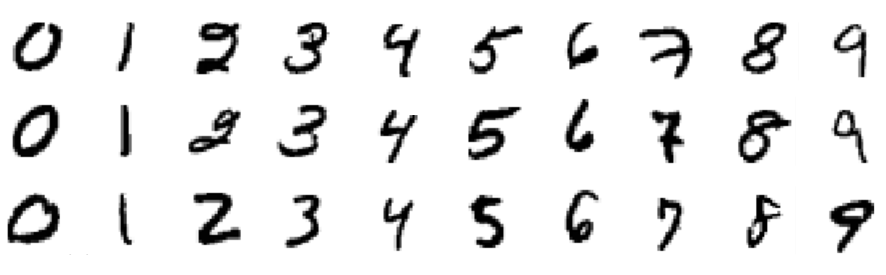Let’s create a Python program to work with this dataset. We will use one file for all of our work in this tutorial. Create a new file called `main.py`:

``````touch main.py

``````

Now open this file in your text editor of choice and add this line of code to the file to import the TensorFlow library:

main.py
``````import tensorflow as tf
``````

Add the following lines of code to your file to import the MNIST dataset and store the image data in the variable `mnist`:

main.py
``````...
from tensorflow.examples.tutorials.mnist import input_data

mnist = input_data.read_data_sets("MNIST_data/", one_hot=True)  # y labels are oh-encoded
``````

When reading in the data, we are using one-hot-encoding to represent the labels (the actual digit drawn, e.g. “3”) of the images. One-hot-encoding uses a vector of binary values to represent numeric or categorical values. As our labels are for the digits 0-9, the vector contains ten values, one for each possible digit. One of these values is set to 1, to represent the digit at that index of the vector, and the rest are set to 0. For example, the digit 3 is represented using the vector `[0, 0, 0, 1, 0, 0, 0, 0, 0, 0]`. As the value at index 3 is stored as 1, the vector therefore represents the digit 3.

To represent the actual images themselves, the 28x28 pixels are flattened into a 1D vector which is 784 pixels in size. Each of the 784 pixels making up the image is stored as a value between 0 and 255. This determines the grayscale of the pixel, as our images are presented in black and white only. So a black pixel is represented by 255, and a white pixel by 0, with the various shades of gray somewhere in between.

We can use the `mnist` variable to find out the size of the dataset we have just imported. Looking at the `num_examples` for each of the three subsets, we can determine that the dataset has been split into 55,000 images for training, 5000 for validation, and 10,000 for testing. Add the following lines to your file:

main.py
``````...
n_train = mnist.train.num_examples  # 55,000
n_validation = mnist.validation.num_examples  # 5000
n_test = mnist.test.num_examples  # 10,000
``````

Now that we have our data imported, it’s time to think about the neural network.

## Step 3 — Defining the Neural Network Architecture

The architecture of the neural network refers to elements such as the number of layers in the network, the number of units in each layer, and how the units are connected between layers. As neural networks are loosely inspired by the workings of the human brain, here the term unit is used to represent what we would biologically think of as a neuron. Like neurons passing signals around the brain, units take some values from previous units as input, perform a computation, and then pass on the new value as output to other units. These units are layered to form the network, starting at a minimum with one layer for inputting values, and one layer to output values. The term hidden layer is used for all of the layers in between the input and output layers, i.e. those “hidden” from the real world.

Different architectures can yield dramatically different results, as the performance can be thought of as a function of the architecture among other things, such as the parameters, the data, and the duration of training.

Add the following lines of code to your file to store the number of units per layer in global variables. This allows us to alter the network architecture in one place, and at the end of the tutorial you can test for yourself how different numbers of layers and units will impact the results of our model:

main.py
``````...
n_input = 784  # input layer (28x28 pixels)
n_hidden1 = 512  # 1st hidden layer
n_hidden2 = 256  # 2nd hidden layer
n_hidden3 = 128  # 3rd hidden layer
n_output = 10  # output layer (0-9 digits)
``````

The following diagram shows a visualization of the architecture we’ve designed, with each layer fully connected to the surrounding layers: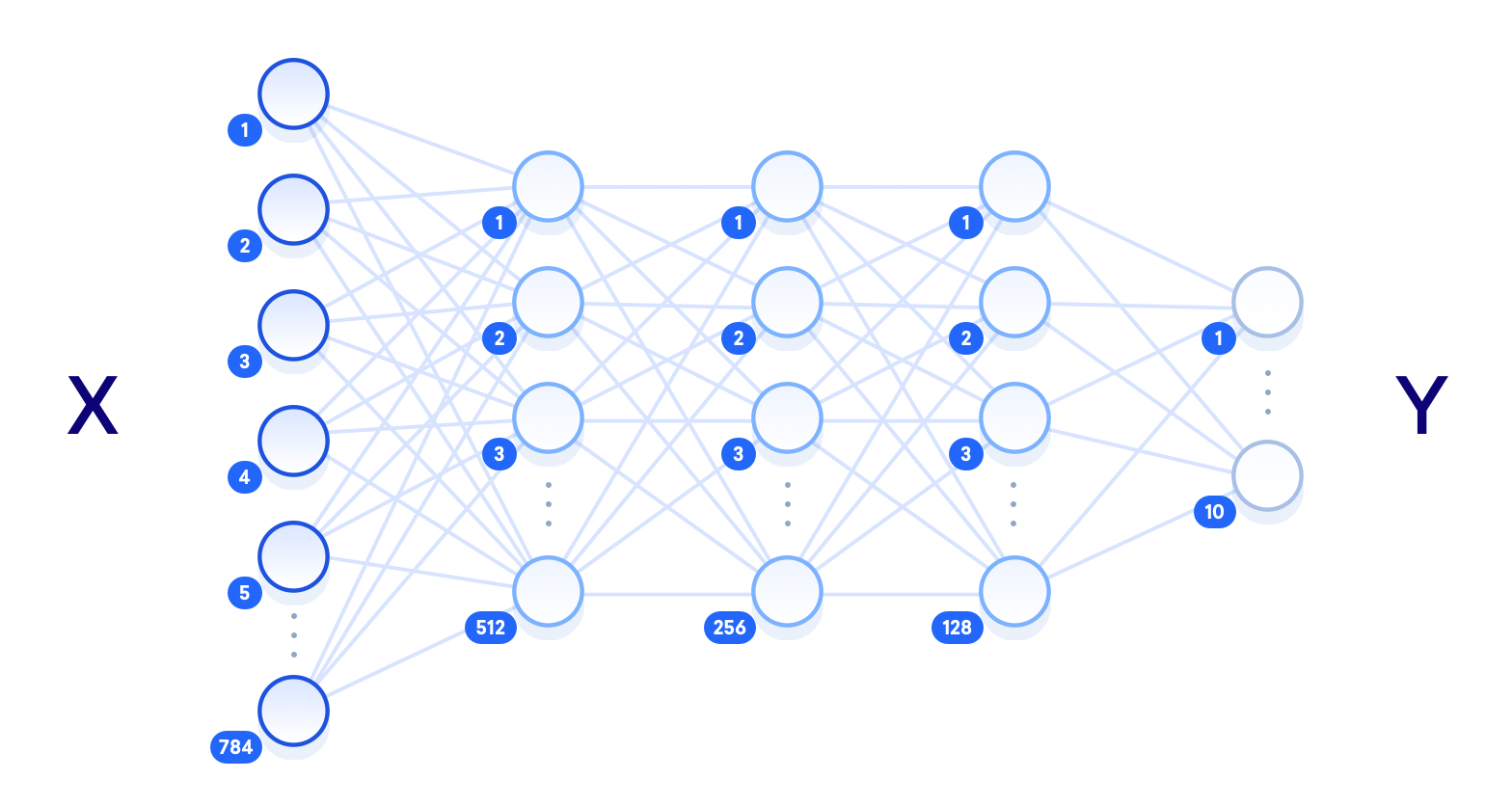The term “deep neural network” relates to the number of hidden layers, with “shallow” usually meaning just one hidden layer, and “deep” referring to multiple hidden layers. Given enough training data, a shallow neural network with a sufficient number of units should theoretically be able to represent any function that a deep neural network can. But it is often more computationally efficient to use a smaller deep neural network to achieve the same task that would require a shallow network with exponentially more hidden units. Shallow neural networks also often encounter overfitting, where the network essentially memorizes the training data that it has seen, and is not able to generalize the knowledge to new data. This is why deep neural networks are more commonly used: the multiple layers between the raw input data and the output label allow the network to learn features at various levels of abstraction, making the network itself better able to generalize.

Other elements of the neural network that need to be defined here are the hyperparameters. Unlike the parameters that will get updated during training, these values are set initially and remain constant throughout the process. In your file, set the following variables and values:

main.py
``````...
learning_rate = 1e-4
n_iterations = 1000
batch_size = 128
dropout = 0.5
``````

The learning rate represents how much the parameters will adjust at each step of the learning process. These adjustments are a key component of training: after each pass through the network we tune the weights slightly to try and reduce the loss. Larger learning rates can converge faster, but also have the potential to overshoot the optimal values as they are updated. The number of iterations refers to how many times we go through the training step, and the batch size refers to how many training examples we are using at each step. The `dropout` variable represents a threshold at which we eliminate some units at random. We will be using `dropout` in our final hidden layer to give each unit a 50% chance of being eliminated at every training step. This helps prevent overfitting.

We have now defined the architecture of our neural network, and the hyperparameters that impact the learning process. The next step is to build the network as a TensorFlow graph.

## Step 4 — Building the TensorFlow Graph

To build our network, we will set up the network as a computational graph for TensorFlow to execute. The core concept of TensorFlow is the tensor, a data structure similar to an array or list. initialized, manipulated as they are passed through the graph, and updated through the learning process.

We’ll start by defining three tensors as placeholders, which are tensors that we’ll feed values into later. Add the following to your file:

main.py
``````...
X = tf.placeholder("float", [None, n_input])
Y = tf.placeholder("float", [None, n_output])
keep_prob = tf.placeholder(tf.float32)
``````

The only parameter that needs to be specified at its declaration is the size of the data we will be feeding in. For `X` we use a shape of `[None, 784]`, where `None` represents any amount, as we will be feeding in an undefined number of 784-pixel images. The shape of `Y` is `[None, 10]` as we will be using it for an undefined number of label outputs, with 10 possible classes. The `keep_prob` tensor is used to control the dropout rate, and we initialize it as a placeholder rather than an immutable variable because we want to use the same tensor both for training (when `dropout` is set to `0.5`) and testing (when `dropout` is set to `1.0`).

The parameters that the network will update in the training process are the `weight` and `bias` values, so for these we need to set an initial value rather than an empty placeholder. These values are essentially where the network does its learning, as they are used in the activation functions of the neurons, representing the strength of the connections between units.

Since the values are optimized during training, we could set them to zero for now. But the initial value actually has a significant impact on the final accuracy of the model. We’ll use random values from a truncated normal distribution for the weights. We want them to be close to zero, so they can adjust in either a positive or negative direction, and slightly different, so they generate different errors. This will ensure that the model learns something useful. Add these lines:

main.py
``````...
weights = {
'w1': tf.Variable(tf.truncated_normal([n_input, n_hidden1], stddev=0.1)),
'w2': tf.Variable(tf.truncated_normal([n_hidden1, n_hidden2], stddev=0.1)),
'w3': tf.Variable(tf.truncated_normal([n_hidden2, n_hidden3], stddev=0.1)),
'out': tf.Variable(tf.truncated_normal([n_hidden3, n_output], stddev=0.1)),
}
``````

For the bias, we use a small constant value to ensure that the tensors activate in the intial stages and therefore contribute to the propagation. The weights and bias tensors are stored in dictionary objects for ease of access. Add this code to your file to define the biases:

main.py
``````...
biases = {
'b1': tf.Variable(tf.constant(0.1, shape=[n_hidden1])),
'b2': tf.Variable(tf.constant(0.1, shape=[n_hidden2])),
'b3': tf.Variable(tf.constant(0.1, shape=[n_hidden3])),
'out': tf.Variable(tf.constant(0.1, shape=[n_output]))
}
``````

Next, set up the layers of the network by defining the operations that will manipulate the tensors. Add these lines to your file:

main.py
``````...
layer_drop = tf.nn.dropout(layer_3, keep_prob)
output_layer = tf.matmul(layer_3, weights['out']) + biases['out']
``````

Each hidden layer will execute matrix multiplication on the previous layer’s outputs and the current layer’s weights, and add the bias to these values. At the last hidden layer, we will apply a dropout operation using our `keep_prob` value of 0.5.

The final step in building the graph is to define the loss function that we want to optimize. A popular choice of loss function in TensorFlow programs is cross-entropy, also known as log-loss, which quantifies the difference between two probability distributions (the predictions and the labels). A perfect classification would result in a cross-entropy of 0, with the loss completely minimized.

We also need to choose the optimization algorithm which will be used to minimize the loss function. A process named gradient descent optimization is a common method for finding the (local) minimum of a function by taking iterative steps along the gradient in a negative (descending) direction. There are several choices of gradient descent optimization algorithms already implemented in TensorFlow, and in this tutorial we will be using the Adam optimizer. This extends upon gradient descent optimization by using momentum to speed up the process through computing an exponentially weighted average of the gradients and using that in the adjustments. Add the following code to your file:

main.py
``````...
cross_entropy = tf.reduce_mean(
tf.nn.softmax_cross_entropy_with_logits(
labels=Y, logits=output_layer
))
``````

We’ve now defined the network and built it out with TensorFlow. The next step is to feed data through the graph to train it, and then test that it has actually learnt something.

## Step 5 — Training and Testing

The training process involves feeding the training dataset through the graph and optimizing the loss function. Every time the network iterates through a batch of more training images, it updates the parameters to reduce the loss in order to more accurately predict the digits shown. The testing process involves running our testing dataset through the trained graph, and keeping track of the number of images that are correctly predicted, so that we can calculate the accuracy.

Before starting the training process, we will define our method of evaluating the accuracy so we can print it out on mini-batches of data while we train. These printed statements will allow us to check that from the first iteration to the last, loss decreases and accuracy increases; they will also allow us to track whether or not we have ran enough iterations to reach a consistent and optimal result:

main.py
``````...
correct_pred = tf.equal(tf.argmax(output_layer, 1), tf.argmax(Y, 1))
accuracy = tf.reduce_mean(tf.cast(correct_pred, tf.float32))
``````

In `correct_pred`, we use the `arg_max` function to compare which images are being predicted correctly by looking at the `output_layer` (predictions) and `Y` (labels), and we use the `equal` function to return this as a list of Booleans. We can then cast this list to floats and calculate the mean to get a total accuracy score.

We are now ready to initialize a session for running the graph. In this session we will feed the network with our training examples, and once trained, we feed the same graph with new test examples to determine the accuracy of the model. Add the following lines of code to your file:

main.py
``````...
init = tf.global_variables_initializer()
sess = tf.Session()
sess.run(init)
``````

The essence of the training process in deep learning is to optimize the loss function. Here we are aiming to minimize the difference between the predicted labels of the images, and the true labels of the images. The process involves four steps which are repeated for a set number of iterations:

• Propagate values forward through the network
• Compute the loss
• Propagate values backward through the network
• Update the parameters

At each training step, the parameters are adjusted slightly to try and reduce the loss for the next step. As the learning progresses, we should see a reduction in loss, and eventually we can stop training and use the network as a model for testing our new data.

Add this code to the file:

main.py
``````...
# train on mini batches
for i in range(n_iterations):
batch_x, batch_y = mnist.train.next_batch(batch_size)
sess.run(train_step, feed_dict={
X: batch_x, Y: batch_y, keep_prob: dropout
})

# print loss and accuracy (per minibatch)
if i % 100 == 0:
minibatch_loss, minibatch_accuracy = sess.run(
[cross_entropy, accuracy],
feed_dict={X: batch_x, Y: batch_y, keep_prob: 1.0}
)
print(
"Iteration",
str(i),
"\t| Loss =",
str(minibatch_loss),
"\t| Accuracy =",
str(minibatch_accuracy)
)
``````

After 100 iterations of each training step in which we feed a mini-batch of images through the network, we print out the loss and accuracy of that batch. Note that we should not be expecting a decreasing loss and increasing accuracy here, as the values are per batch, not for the entire model. We use mini-batches of images rather than feeding them through individually to speed up the training process and allow the network to see a number of different examples before updating the parameters.

Once the training is complete, we can run the session on the test images. This time we are using a `keep_prob` dropout rate of `1.0` to ensure all units are active in the testing process.

Add this code to the file:

main.py
``````...
test_accuracy = sess.run(accuracy, feed_dict={X: mnist.test.images, Y: mnist.test.labels, keep_prob: 1.0})
print("\nAccuracy on test set:", test_accuracy)
``````

It’s now time to run our program and see how accurately our neural network can recognize these handwritten digits. Save the `main.py` file and execute the following command in the terminal to run the script:

``````python main.py

``````

You’ll see an output similar to the following, although individual loss and accuracy results may vary slightly:

``````OutputIteration 0 	| Loss = 3.67079 	| Accuracy = 0.140625
Iteration 100 	| Loss = 0.492122 	| Accuracy = 0.84375
Iteration 200 	| Loss = 0.421595 	| Accuracy = 0.882812
Iteration 300 	| Loss = 0.307726 	| Accuracy = 0.921875
Iteration 400 	| Loss = 0.392948 	| Accuracy = 0.882812
Iteration 500 	| Loss = 0.371461 	| Accuracy = 0.90625
Iteration 600 	| Loss = 0.378425 	| Accuracy = 0.882812
Iteration 700 	| Loss = 0.338605 	| Accuracy = 0.914062
Iteration 800 	| Loss = 0.379697 	| Accuracy = 0.875
Iteration 900 	| Loss = 0.444303 	| Accuracy = 0.90625

Accuracy on test set: 0.9206
``````

To try and improve the accuracy of our model, or to learn more about the impact of tuning hyperparameters, we can test the effect of changing the learning rate, the dropout threshold, the batch size, and the number of iterations. We can also change the number of units in our hidden layers, and change the amount of hidden layers themselves, to see how different architectures increase or decrease the model accuracy.

To demonstrate that the network is actually recognizing the hand-drawn images, let’s test it on a single image of our own.

If you are on a local machine and you would like to use your own hand-drawn number, you can use a graphics editor to create your own 28x28 pixel image of a digit. Otherwise, you can use `curl` to download the following sample test image to your server or computer:

``````curl -O https://raw.githubusercontent.com/do-community/tensorflow-digit-recognition/master/test_img.png

``````

Open the `main.py` file in your editor and add the following lines of code to the top of the file to import two libraries necessary for image manipulation.

main.py
``````import numpy as np
from PIL import Image
...
``````

Then at the end of the file, add the following line of code to load the test image of the handwritten digit:

main.py
``````...
img = np.invert(Image.open("test_img.png").convert('L')).ravel()
``````

The `open` function of the `Image` library loads the test image as a 4D array containing the three RGB color channels and the Alpha transparency. This is not the same representation we used previously when reading in the dataset with TensorFlow, so we’ll need to do some extra work to match the format.

First, we use the `convert` function with the `L` parameter to reduce the 4D RGBA representation to one grayscale color channel. We store this as a `numpy` array and invert it using `np.invert`, because the current matrix represents black as 0 and white as 255, whereas we need the opposite. Finally, we call `ravel` to flatten the array.

Now that the image data is structured correctly, we can run a session in the same way as previously, but this time only feeding in the single image for testing.

Add the following code to your file to test the image and print the outputted label.

main.py
``````...
prediction = sess.run(tf.argmax(output_layer, 1), feed_dict={X: [img]})
print ("Prediction for test image:", np.squeeze(prediction))
``````

The `np.squeeze` function is called on the prediction to return the single integer from the array (i.e. to go from  to 2). The resulting output demonstrates that the network has recognized this image as the digit 2.

``````OutputPrediction for test image: 2
``````

You can try testing the network with more complex images –– digits that look like other digits, for example, or digits that have been drawn poorly or incorrectly –– to see how well it fares.

## Conclusion

In this tutorial you successfully trained a neural network to classify the MNIST dataset with around 92% accuracy and tested it on an image of your own. Current state-of-the-art research achieves around 99% on this same problem, using more complex network architectures involving convolutional layers. These use the 2D structure of the image to better represent the contents, unlike our method which flattened all the pixels into one vector of 784 units. You can read more about this topic on the TensorFlow website, and see the research papers detailing the most accurate results on the MNIST website.

Now that you know how to build and train a neural network, you can try and use this implementation on your own data, or test it on other popular datasets such as the Google StreetView House Numbers, or the CIFAR-10 dataset for more general image recognition.

]]>
Ellie Birbeck 2021-08-11T20:37:47.451Z
<![CDATA[Best way to deploy react/django web app on digital ocean]]> tag:www.digitalocean.com,2005:/community/questions/best-way-to-deploy-react-django-web-app-on-digital-ocean 2021-05-11T07:58:06.162Z I am building web application with django as a backend and reactJS as a frontend. I need to know which hosting solution works best for me ? What I am looking for:

1. I need to store a lot of images so I need a lot of disk space (my estimate around 500GB at a moment)
2. Currently, there would only be 50 users of my app using my website 24/7 so right now I may not need huge amount of computing power but I may be doing some face recognition or detection on around 10,000 per day.
3. Also, I am not sure how this django + react app works with deployment so if you can guide me ? like cloud hosting or linux virtual machine? I have no idea about it which is best in terms of performance+pricing

]]>
haiderabbas1982000 2021-05-11T07:58:06.162Z
<![CDATA[How To Install and Use PyTorch]]> tag:www.digitalocean.com,2005:/community/tutorials/how-to-install-and-use-pytorch 2021-02-11T16:11:04.170Z The author selected the International Medical Corps to receive a donation as part of the Write for DOnations program.

### Introduction

PyTorch is a framework developed by Facebook AI Research for deep learning, featuring both beginner-friendly debugging tools and a high-level of customization for advanced users, with researchers and practitioners using it across companies like Facebook and Tesla. Applications include computer vision, natural language processing, cryptography, and more. Whereas more advanced DigitalOcean articles, like Introduction to PyTorch, discuss how PyTorch works and what you can build with PyTorch, this tutorial will focus on installing PyTorch.

In this tutorial, you’ll install PyTorch’s “CPU support only” version in three steps. This installation is ideal for people looking to install and use PyTorch but who don’t have an Nvidia graphics card. In particular, you’ll install PyTorch in a Python virtual environment with virtualenv. This approach isolates the PyTorch installation, allowing you to install different PyTorch versions for each project. Once you complete the installation, you’ll validate your installation by running a short PyTorch program and then use PyTorch to perform image classification.

While you won’t need prior experience in practical deep learning or PyTorch to follow along with this tutorial, we’ll assume some familiarity with machine learning terms and concepts such as tensors. You can learn more about these concepts in An Introduction to Machine Learning.

## Prerequisites

To complete this tutorial, you will need a local development environment for Python 3 with at least 1GB of RAM. You can follow How to Install and Set Up a Local Programming Environment for Python 3 to set up Python and the essentials for your programming environment.

## Step 1 — Installing PyTorch

Let’s create a workspace for this project and install the dependencies you’ll need. You’ll call your workspace `pytorch`:

``````mkdir ~/pytorch

``````

Make a directory to hold all your assets:

``````mkdir ~/pytorch/assets

``````

Navigate to the `pytorch` directory:

``````cd ~/pytorch

``````

Then create a new virtual environment for the project:

``````python3 -m venv pytorch

``````

``````source pytorch/bin/activate

``````

Then install PyTorch. On macOS, install PyTorch with the following command:

``````pip install torch torchvision

``````

On Linux and Windows, use the following commands for a CPU-only build:

``````pip install torch==1.7.1+cpu torchvision==0.8.2+cpu -f https://download.pytorch.org/whl/torch_stable.html

``````

Notice that you have also included `torchvision` by default. This sub-library includes several utilities specific to computer vision, which you’ll use later on in this tutorial. You’ll then receive output confirming PyTorch’s installation:

``````OutputCollecting torch
|████████████████████████████████| 108.9 MB 8.3 MB/s

. . .

Successfully installed pillow-8.1.0 torch-1.7.1 torchvision-0.8.2
``````

Note: If you’d like to deactivate your virtual environment at any time, the command is:

``````deactivate

``````

To reactivate the environment later, navigate to your project directory and run `source pytorch/bin/activate`.

Now, that you have installed PyTorch, you’ll make sure the PyTorch installation works.

## Step 2 — Validating Installation

To validate the installation of PyTorch, you’re going to run a small program in PyTorch as a non-root user. Rather than creating a Python file, you’ll create this program using Python’s interactive console.

To write the program, start up your Python interpreter with the following command:

``````python3

``````

``````

``````

This is the prompt for the Python interpreter, and it indicates that it’s ready for you to start entering some Python statements.

First, type this line to import the PyTorch package. Press `ENTER` after typing in the line of code:

``````import torch

``````

Define a vector of zeros. For now, think of a vector as a collection of numbers, or specifically, a list of numbers. In more detail: A vector is an “arrow” in space, indicating both direction (where the arrow points), and magnitude (how long the arrow is). Next, you create a vector using a list of three numbers with `Tensor()`. This is a three-dimensional vector, which is an arrow in three-dimensional space.

Run the following Python:

``````torch.Tensor([0, 0, 0])

``````

``````Outputtensor([0., 0., 0.])
``````

This indicates the PyTorch installation was successful. Exit the Python interactive console by pressing `CTRL+D`. Next, you will build an image classifier using PyTorch.

## Step 3 — Using PyTorch for Image Classification

Now that you’ve validated the PyTorch installation, you will set up an image classifier.

An image classifier accepts images as input and outputs a predicted class (like `Cat` or `Dog`). Image classifiers are the conventional “Hello World” equivalent in deep learning frameworks. Besides convention, there are a few great reasons to start with image classification. First, many image classifiers can produce predictions on a commodity CPU, without needing extensive GPU resources. Second, it is straightforward to know that your image classifier is working (or not), based on the predicted class. This is less true of other neural networks that, for example, generate text.

In this tutorial, you will use image classifiers that have already been trained. We call these pretrained image classifiers. In particular, you will use image classifiers to predict the class of an image. Prediction is alternatively called inference. In short, you will be running inference on a pretrained image classifier.

``````wget -O ~/pytorch/assets/imagenet_idx_to_label.json https://raw.githubusercontent.com/do-community/tricking-neural-networks/master/utils/imagenet_idx_to_label.json

``````

Download the following Python script, which will load an image, load a neural network with its weights, and classify the image using the neural network:

``````wget https://raw.githubusercontent.com/do-community/tricking-neural-networks/master/step_2_pretrained.py

``````

Note: For a more detailed walkthrough of this file `step_2_pretrained.py`, please read Step 2 — Running a Pretrained Animal Classifier in the How To Trick a Neural Network tutorial.

Download the following image of a dog to run the image classifier on.Use the following command to do so:

``````wget -O assets/dog.jpg https://assets.digitalocean.com/articles/trick_neural_network/step2a.png

``````

Finally, run the pretrained image classifier on the newly downloaded image. Pretrained means this model has already been trained and will be able to predict classes, accurately, straightaway:

``````python step_2_pretrained.py assets/dog.jpg

``````

This will produce the following output, showing your animal classifier works as expected:

``````OutputPrediction: Pembroke, Pembroke Welsh corgi
``````

That concludes running inference with your pretrained model. If you’d like to use another image, you can do this by changing the first argument to your `python3 step_2_pretrained.py` command. For the argument, you’d pass in the relative path of the image file. In the next step, we’ll summarize a few recommended tools for working in PyTorch.

## The PyTorch Ecosystem

In this section, we recommend several frameworks and libraries to get started with, as you work with PyTorch. Although these additional libraries may not be beneficial until later in your PyTorch journey, knowing that these tools exist is useful, so you can leverage them when you are ready.

For each deep learning library, there is a canonical higher-level framework to use. PyTorch was the exception to this rule for its first few years. However, Facebook AI Research released two frameworks in 2019 that have grown quickly in popularity. Note that neither library is fully mature as of February 2021, and as a result, native PyTorch tutorials are a much more friendly place to start.

There are several deep-learning-library-agnostic tools that are additionally useful to integrate as well. Both of these are prolific in both the research and industry communities.

• Weights and Biases is designed for experiment tracking, particularly useful for a) communicating between collaborators, b) keeping experiments organized, and c) quickly visualizing many experimental results simultaneously.
• Tensorboard is ostensibly designed for Tensorflow. However, because PyTorch does not natively support a visualization dashboard, PyTorch practitioners adopted Tensorboard. Find the PyTorch tutorial for Tensorboard visualizations on the PyTorch website.

To deploy a PyTorch model to production, there are several commonly used options:

• TorchScript is the natively supported deployment option for PyTorch models. However, most model deployment in 2021 uses one of the two following methods.
• ONNX is an open-source format for all deep learning frameworks, supporting not just deployment but interoperability between deep learning frameworks. In practice, converting from a PyTorch model to the ONNX format takes some debugging to make work. This has seen incremental improvement over the years, as PyTorch increases native support for ONNX exports.
• Caffe2 is the most commonly used deployment option of these three. Although Caffe2 was itself a standalone deep learning framework, it was subsequently merged with PyTorch in 2018. You can learn more about deploying with Caffe2 on the PyTorch tutorial website.

There are also several application-focused PyTorch GitHub repositories, useful for reproducing and then building off of state-of-the-art results in their respective areas of research:

• Detectron2 for computer vision supports object detection, object segmentation, keypoint estimation, and more. It also includes utilities for exporting a model in either Torchscript or Caffe2.
• Transformers for natural language processing supports a large number of state-of-the-art models and their associated tokenizers.

You can find more PyTorch libraries in the PyTorch Ecosystem.

## Conclusion

You’ve installed PyTorch in a Python virtual environment and validated that PyTorch works by running a couple of examples. You now have the tools to explore machine learning further. For your next step in developing deep learning know-how, check out an Introduction to PyTorch: Build a Neural Network to Recognize Handwritten Digits. We’ll cover more than just PyTorch; we’ll also discuss the foundations of machine learning.

To learn more about PyTorch, see the official tutorials. You can also get started with other popular deep learning frameworks, including Tensorflow. For more machine learning content, check out our Machine Learning topic page.

]]>
Alvin Wan 2021-02-11T16:11:04.170Z
<![CDATA[Introduction to PyTorch: Build a Neural Network to Recognize Handwritten Digits]]> tag:www.digitalocean.com,2005:/community/tutorials/introduction-to-pytorch-build-a-neural-network-to-recognize-handwritten-digits 2021-01-20T16:06:05.799Z The author selected the Code 2040 to receive a donation as part of the Write for DOnations program.

### Introduction

Machine learning is a field of computer science that finds patterns in data. As of 2021, machine learning practitioners use these patterns to detect lanes for self-driving cars; train a robot hand to solve a Rubik’s cube; or generate images of dubious artistic taste. As machine learning models grow more accurate and performant, we see increasing adoption in mainstream applications and products.

Deep learning is a subset of machine learning that focuses on particularly complex models, termed neural networks. In later, advanced DigitalOcean articles (like this tutorial on building an Atari bot), we will formally define what “complex” means. Neural networks are the highly accurate and hype-inducing modern-day models your hear about, with applications across a wide range of tasks. In this tutorial, you will focus on one specific task called object recognition, or image classification. Given an image of a handwritten digit, your model will predict which digit is shown.

You will build, train, and evaluate deep neural networks in PyTorch, a framework developed by Facebook AI Research for deep learning. When compared to other deep learning frameworks, like Tensorflow, PyTorch is a beginner-friendly framework with debugging features that aid in the building process. It’s also highly customizable for advanced users, with researchers and practitioners using it across companies like Facebook and Tesla. By the end of this tutorial, you will be able to:

• Build, train, and evaluate a deep neural network in PyTorch
• Understand the risks of applying deep learning

While you won’t need prior experience in practical deep learning or PyTorch to follow along with this tutorial, we’ll assume some familiarity with machine learning terms and concepts such as training and testing, features and labels, optimization, and evaluation. You can learn more about these concepts in An Introduction to Machine Learning.

## Prerequisites

To complete this tutorial, you will need a local development environment for Python 3 with at least 1GB of RAM. You can follow How to Install and Set Up a Local Programming Environment for Python 3 to configure everything you need.

## Step 1 — Creating Your Project and Installing Dependencies

Let’s create a workspace for this project and install the dependencies you’ll need. You’ll call your workspace `pytorch`:

``````mkdir ~/pytorch

``````

Navigate to the `pytorch` directory:

``````cd ~/pytorch

``````

Then create a new virtual environment for the project:

``````python3 -m venv pytorch

``````

``````source pytorch/bin/activate

``````

Then install PyTorch. On macOS, install PyTorch with the following command:

``````python -m pip install torch==1.4.0 torchvision==0.5.0

``````

On Linux and Windows, use the following commands for a CPU-only build:

``````pip install torch==1.4.0+cpu torchvision==0.5.0+cpu -f https://download.pytorch.org/whl/torch_stable.html
pip install torchvision

``````

With the dependencies installed, you will now build your first neural network.

## Step 2 — Building a “Hello World” Neural Network

In this step, you will build your first neural network and train it. You will learn about two sub-libraries in Pytorch, `torch.nn` for neural network operations and `torch.optim` for neural network optimizers. To understand what an “optimizer” is, you will also learn about an algorithm called gradient descent. Throughout this tutorial, you will use the following five steps to build and train models:

1. Build a computation graph
2. Set up optimizers
3. Set up criterion
4. Set up data
5. Train the model

In this first section of the tutorial, you will build a small model with a manageable dataset. Start by creating a new file `step_2_helloworld.py`, using `nano` or your favorite text editor:

``````nano step_2_helloworld.py

``````

You will now write a short 18-line snippet that trains a small model. Start by importing several PyTorch utilities:

step_2_helloworld.py
``````import torch
import torch.nn as nn
import torch.optim as optim
``````

Here, you alias PyTorch libraries to several commonly used shortcuts:

• `torch` contains all PyTorch utilities. However, routine PyTorch code includes a few extra imports. We follow the same convention here, so that you can understand PyTorch tutorials and random code snippets online.
• `torch.nn` contains utilities for constructing neural networks. This is often denoted `nn`.
• `torch.optim` contains training utilities. This is often denoted `optim`.

Next, define the neural network, training utilities, and the dataset:

step_2_helloworld.py
``````. . .
net = nn.Linear(1, 1)  # 1. Build a computation graph (a line!)
optimizer = optim.SGD(net.parameters(), lr=0.1)  # 2. Setup optimizers
criterion = nn.MSELoss()  # 3. Setup criterion
x, target = torch.randn((1,)), torch.tensor([0.])  # 4. Setup data
. . .
``````

Here, you define several necessary parts of any deep learning training script:

• `net = ...` defines the “neural network”. In this case, the model is a line of the form `y = m * x`; the parameter `nn.Linear(1, 1)` is the slope of your line. This model parameter `nn.Linear(1, 1)` will be updated during training. Note that `torch.nn` (aliased with `nn`) includes many deep learning operations, like the fully connected layers used here (`nn.Linear`) and convolutional layers (`nn.Conv2d`).
• `optimizer = ...` defines the optimizer. This optimizer determines how the neural network will learn. We will discuss optimizers in more detail after writing a few more lines of code. Note that `torch.optim` (aliased to `optim`) includes many such optimizers that you can use.
• `criterion = ...` defines the loss. In short, the loss defines what your model is trying to minimize. For your basic model of a line, the goal is to minimize the difference between your line’s predicted y-values and the actual y-values in the training set. Note that `torch.nn` (aliased with `nn`) includes many other loss functions you can use.
• `x, target = ...` defines your “dataset”. Right now, the dataset is just one coordinate—one x value and one y value. In this case, the `torch` package itself offers `tensor`, to create a new tensor, and `randn` to create a tensor with random values.

Finally, train the model by iterating over the dataset ten times. Each time, you adjust the model’s parameter:

step_2_helloworld.py
``````. . .
# 5. Train the model
for i in range(10):
output = net(x)
loss = criterion(output, target)
print(round(loss.item(), 2))

loss.backward()
optimizer.step()
``````

Your general goal is to minimize the loss, by adjusting the slope of the line. To effect this, this training code implements an algorithm called gradient descent. The intuition for gradient descent is as follows: Imagine you’re looking straight down at a bowl. The bowl has many points on it, and each point corresponds to a different parameter value. The bowl itself is the loss surface: the center of the bowl—the lowest point—indicates the best model with the lowest loss. This is the optimum. The fringes of the bowl—the highest points, and the parts of the bowl closest to you—hold the worst models with the highest loss.

To find the best model with the lowest loss:

1. With `net = nn.Linear(1, 1)` you initialize a random model. This is equivalent to picking a random point on the bowl.
2. In the `for i in range(10)` loop, you begin training. This is equivalent to stepping closer to the center of the bowl.
3. The direction of each step is given by the gradient. You will skip a formal proof here, but in summary, the negative gradient points to the lowest point in the bowl.
4. With `lr=0.1` in `optimizer = ...`, you specify the step size. This determines how large each step can be.

In just ten steps, you reach the center of the bowl, the best possible model with the lowest possible loss. For a visualization of gradient descent, see Distill’s “Why Momentum Really Works,” first figure at the top of the page.

The last three lines of this code are also important:

• `net.zero_grad` clears all gradients that may have been leftover from the previous step iterate.
• `loss.backward` computes new gradients.
• `optimizer.step` uses those gradients to take steps. Notice that you didn’t compute gradients yourself. This is because PyTorch, and other deep learning libraries like it, automatically differentiate.

This now concludes your “hello world” neural network. Save and close your file.

Double-check that your script matches `step_2_helloworld.py`. Then, run the script:

``````python step_2_helloworld.py

``````

Your script will output the following:

``````Output0.33
0.19
0.11
0.07
0.04
0.02
0.01
0.01
0.0
0.0
``````

Notice that your loss continually decreases, showing that your model is learning. There are two other implementation details to note, when using PyTorch:

1. PyTorch uses `torch.Tensor` to hold all data and parameters. Here, `torch.randn` generates a tensor with random values, with the provided shape. For example, a `torch.randn((1, 2))` creates a 1x2 tensor, or a 2-dimensional row vector.
2. PyTorch supports a wide variety of optimizers. This features `torch.optim.SGD`, otherwise known as stochastic gradient descent (SGD). Roughly speaking, this is the algorithm described in this tutorial, where you took steps toward the optimum. There are more-involved optimizers that add extra features on top of SGD. There are also many losses, with `torch.nn.MSELoss` being just one of them.

This concludes your very first model on a toy dataset. In the next step, you will replace this small model with a neural network and the toy dataset with a commonly used machine learning benchmark.

## Step 3 — Training Your Neural Network on Handwritten Digits

In the previous section, you built a small PyTorch model. However, to better understand the benefits of PyTorch, you will now build a deep neural network using `torch.nn.functional`, which contains more neural network operations, and `torchvision.datasets`, which supports many datasets you can use, out of the box. In this section, you will build a relatively complex, custom model with a premade dataset.

You’ll use convolutions, which are pattern-finders. For images, convolutions look for 2D patterns at various levels of “meaning”: Convolutions directly applied to the image are looking for “lower-level” features such as edges. However, convolutions applied to the outputs of many other operations may be looking for “higher-level” features, such as a door. For visualizations and a more thorough walkthrough of convolutions, see part of Stanford’s deep learning course.

You will now expand on the first PyTorch model you built, by defining a slightly more complex model. Your neural network will now contain two convolutions and one fully connected layer, to handle image inputs.

Start by creating a new file `step_3_mnist.py`, using your text editor:

``````nano step_3_mnist.py

``````

You will follow the same five-step algorithm as before:

1. Build a computation graph
2. Set up optimizers
3. Set up criterion
4. Set up data
5. Train the model

First, define your deep neural network. Note this is a pared down version of other neural networks you may find on MNIST—this is intentional, so that you can train your neural network on your laptop:

step_3_mnist.py
``````import torch
import torch.nn as nn
import torch.optim as optim
import torch.nn.functional as F

from torchvision import datasets, transforms
from torch.optim.lr_scheduler import StepLR

# 1. Build a computation graph
class Net(nn.Module):
def __init__(self):
super(Net, self).__init__()
self.conv1 = nn.Conv2d(1, 32, 3, 1)
self.conv2 = nn.Conv2d(32, 64, 3, 1)
self.fc = nn.Linear(1024, 10)

def forward(self, x):
x = F.relu(self.conv1(x))
x = F.relu(self.conv2(x))
x = F.max_pool2d(x, 1)
x = torch.flatten(x, 1)
x = self.fc(x)
output = F.log_softmax(x, dim=1)
return output
net = Net()
. . .
``````

Here, you define a neural network class, inheriting from `nn.Module`. All operations in the neural network (including the neural network itself) must inherit from `nn.Module`. The typical paradigm, for your neural network class, is as follows:

1. In the constructor, define any operations needed for your network. In this case, you have two convolutions and a fully connected layer. (A tip to remember: The constructor always starts with `super().__init__()`.) PyTorch expects the parent class to be initialized before assigning modules (for example, `nn.Conv2d`) to instance attributes (`self.conv1`).
2. In the `forward` method, run the initialized operations. This method determines the neural network architecture, explicitly defining how the neural network will compute its predictions.

This neural network uses a few different operations:

• `nn.Conv2d`: A convolution. Convolutions look for patterns in the image. Earlier convolutions look for “low-level” patterns like edges. Later convolutions in the network look for “high-level” patterns like legs on a dog, or ears.
• `nn.Linear`: A fully connected layer. Fully connected layers relate all input features to all output dimensions.
• `F.relu`, `F.max_pool2d`: These are types of non-linearities. (A non-linearity is any function that is not linear.) `relu` is the function `f(x) = max(x, 0)`. `max_pool` takes the maximum value in every patch of values. In this case, you take the maximum value across the entire image.
• `log_softmax`: normalizes all of the values in a vector, so that the values sum to 1.

Second, like before, define the optimizer. This time, you will use a different optimizer and a different hyper-parameter setting. Hyper-parameters configure training, whereas training adjusts model parameters. These hyper-parameter settings are taken from the PyTorch MNIST example:

step_3_mnist.py
``````. . .
. . .
``````

Third, unlike before, you will now use a different loss. This loss is used for classification problems, where the output of your model is a class index. In this particular example, the model will output the digit (possibly any number from 0 to 9) contained in the input image:

step_3_mnist.py
``````. . .
criterion = nn.NLLLoss()  # 3. Setup criterion
. . .
``````

Fourth, set up the data. In this case, you will set up a dataset called MNIST, which features handwritten digits. Deep Learning 101 tutorials often use this dataset. Each image is a small 28x28 px image containing a handwritten digit, and the goal is to classify each handwritten digit as 0, 1, 2, … or 9:

step_3_mnist.py
``````. . .
# 4. Setup data
transform = transforms.Compose([
transforms.Resize((8, 8)),
transforms.ToTensor(),
transforms.Normalize((0.1307,), (0.3081,))
])
train_dataset = datasets.MNIST(
. . .
``````

Here, you preprocess the images in `transform = ...` by resizing the image, converting the image to a PyTorch tensor, and normalizing the tensor to have mean 0 and variance 1.

In the next two lines, you set `train=True`, as this is the training dataset and `download=True` so that you download the dataset if it is not already.

`batch_size=512` determines how many images the network is trained on, at once. Barring ridiculously large batch sizes (for example, tens of thousands), larger batches are preferable for roughly faster training.

Fifth, train the model. In the following code block, you make minimal modifications. Instead of running ten times on the same sample, you will now iterate over all samples in the provided dataset once. By passing over all samples once, the following is training for one epoch:

step_3_mnist.py
``````. . .
# 5. Train the model
output = net(inputs)
loss = criterion(output, target)
print(round(loss.item(), 2))

loss.backward()
optimizer.step()
. . .
``````

Double-check that your script matches `step_3_mnist.py`. Then, run the script.

``````python step_3_mnist.py

``````

Your script will output the following:

``````Output2.31
2.18
2.03
1.78
1.52
1.35
1.3
1.35
1.07
1.0
...
0.21
0.2
0.23
0.12
0.12
0.12
``````

Notice that the final loss is less than 10% of the initial loss value. This means that your neural network is training correctly.

That concludes training. However, the loss of 0.12 is difficult to reason about: we don’t know if 0.12 is “good” or “bad”. To assess how well your model is performing, you next compute an accuracy for this classification model.

## Step 4 — Evaluating Your Neural Network

Earlier, you computed loss values on the train split of your dataset. However, it is good practice to keep a separate validation split of your dataset. You use this validation split to compute the accuracy of your model. However, you can’t use it for training. Following, you set up the validation dataset and evaluate your model on it. In this step, you will use the same PyTorch utilities from before, including `torchvision.datasets` for the MNIST dataset.

Start by copying your `step_3_mnist.py` file into `step_4_eval.py`. Then, open the file:

``````cp step_3_mnist.py step_4_eval.py
nano step_4_eval.py

``````

First, set up the validation dataset:

step_4_eval.py
``````. . .
val_dataset = datasets.MNIST(
. . .
``````

At the end of your file, after the training loop, add a validation loop:

step_4_eval.py
``````    . . .
optimizer.step()

correct = 0.
net.eval()
output = net(inputs)
_, pred = output.max(1)
correct += (pred == target).sum()
accuracy = correct / len(val_dataset) * 100.
print(f'{accuracy:.2f}% correct')
``````

Here, the validation loop performs a few operations to compute accuracy:

• Running `net.eval()` ensures that your neural network is in evaluation mode and ready for validation. Several operations are run differently in evaluation mode than when in training mode.
• Iterating over all inputs and labels in `val_loader`.
• Running the model `net(inputs)` to obtain probabilities for each class.
• Finding the class with the highest probability `output.max(1)`. `output` is a tensor with dimensions `(n, k)` for `n` samples and `k` classes. The `1` means you compute the max along the index `1` dimension.
• Computing the number of images that were classified correctly: `pred == target` computes a boolean-valued vector. `.sum()` casts these booleans to integers and effectively computes the number of true values.
• `correct / len(val_dataset)` finally computes the percent of images classified correctly.

Double-check that your script matches `step_4_eval.py`. Then, run the script:

``````python step_4_eval.py

``````

Your script will output the following. Note the specific loss values and final accuracy may vary:

``````Output2.31
2.21
...
0.14
0.2
89% correct
``````

You have now trained your very first deep neural network. You can make further modifications and improvements by tuning hyper-parameters for training: This includes different numbers of epochs, learning rates, and different optimizers. We include a sample script with tuned hyper-parameters; this script trains the same neural network but for 10 epochs, obtaining 97% accuracy.

## Risks of Deep Learning

One gotcha is that deep learning does not always obtain state-of-the-art results. Deep learning works well in feature-rich, data-rich scenarios but conversely performs poorly in data-sparse, feature-sparse regimes. Whereas there is active research in deep learning’s weak areas, many other machine learning techniques are already well-suited for feature-sparse regimes, such as decision trees, linear regression, or support vector machines (SVM).

Another gotcha is that deep learning is not well understood. There are no guarantees for accuracy, optimality, or even convergence. On the other hand, classic machine learning techniques are well-studied and are relatively interpretable. Again, there is active research to address this lack of interpretability in deep learning. You can read more in “What Explainable AI fails to explain (and how we fix that)”.

Most importantly, lack of interpretability in deep learning leads to overlooked biases. For example, researchers from UC Berkeley were able to show a model’s gender bias in captioning (“Women also Snowboard”). Other research efforts focus on societal issues such as “Fairness” in machine learning. Given these issues are undergoing active research, it is difficult to recommend a prescribed diagnosis for biases in models. As a result, it is up to you, the practitioner, to apply deep learning responsibly.

## Conclusion

PyTorch is deep learning framework for enthusiasts and researchers alike. To get acquainted with PyTorch, you have both trained a deep neural network and also learned several tips and tricks for customizing deep learning.

You can also use a pre-built neural network architecture instead of building your own. Here is a link to an optional section: Use Existing Neural Network Architecture on Google Colab that you can try. For demonstration purposes, this optional step trains a much larger model with much larger images.

Check out our other articles to dive deeper into machine learning and related fields:

• Is your model complex enough? Too complex? Learn about the bias-variance tradeoff in Bias-Variance for Deep Reinforcement Learning: How To Build a Bot for Atari with OpenAI Gym to find out. In this article, we build AI bots for Atari Games and explore a field of research called Reinforcement Learning. Alternatively, find a visual explanation of the bias-variance trade-off in this Understanding the Bias-Variance Trade-off article.
• How does a machine learning model process images? Learn more in Build an Emotion-Based Dog Filter. In this article, we discuss how models process and classify images, in more detail, exploring a field of research called Computer Vision.
• Can a neural network be fooled? Learn how to in Tricking a Neural Network. In this article, we explore adversarial machine learning, a field of research that devises both attacks and defenses for neural networks for more robust real-world deep learning deployments.
• How can we better understand how neural networks work? Read one class of approaches called “Explainable AI” in How To Visualize and Interpret Neural Networks. In this article, we explore explainable AI, and in particular visualize pixels that the neural networks believes are important for its predictions.
]]>
Alvin Wan 2021-01-20T16:06:05.799Z
<![CDATA[How To Visualize and Interpret Neural Networks in Python]]> tag:www.digitalocean.com,2005:/community/tutorials/how-to-visualize-and-interpret-neural-networks 2020-11-23T19:21:07.559Z The author selected Open Sourcing Mental Illness to receive a donation as part of the Write for DOnations program.

### Introduction

Neural networks achieve state-of-the-art accuracy in many fields such as computer vision, natural-language processing, and reinforcement learning. However, neural networks are complex, easily containing hundreds of thousands, or even, millions of operations (MFLOPs or GFLOPs). This complexity makes interpreting a neural network difficult. For example: How did the network arrive at the final prediction? Which parts of the input influenced the prediction? This lack of understanding is exacerbated for high-dimensional inputs like images: What does an explanation for an image classification even look like?

Research in Explainable AI (XAI) works to answers these questions with a number of different explanations. In this tutorial, you’ll specifically explore two types of explanations: 1. Saliency maps, which highlight the most important parts of the input image; and 2. decision trees, which break down each prediction into a sequence of intermediate decisions. For both of these approaches, you’ll produce code that generates these explanations from a neural network.

Along the way, you’ll also use deep-learning Python library `PyTorch`, computer-vision library `OpenCV`, and linear-algebra library `numpy`. By following this tutorial, you will gain an understanding of current XAI efforts to understand and visualize neural networks.

## Prerequisites

To complete this tutorial, you will need the following:

You can find all the code and assets from this tutorial in this repository.

## Step 1 — Creating Your Project and Installing Dependencies

Let’s create a workspace for this project and install the dependencies you’ll need. You’ll call your workspace `XAI`, short for `Explainable Artificial Intelligence`:

``````mkdir ~/XAI

``````

Navigate to the `XAI` directory:

``````cd ~/XAI

``````

Make a directory to hold all your assets:

``````mkdir ~/XAI/assets

``````

Then create a new virtual environment for the project:

``````python3 -m venv xai

``````

``````source xai/bin/activate

``````

Then install PyTorch, a deep-learning framework for Python that you’ll use in this tutorial.

On macOS, install PyTorch with the following command:

``````python -m pip install torch==1.4.0 torchvision==0.5.0

``````

On Linux and Windows, use the following commands for a CPU-only build:

``````pip install torch==1.4.0+cpu torchvision==0.5.0+cpu -f https://download.pytorch.org/whl/torch_stable.html
pip install torchvision

``````

Now install prepackaged binaries for `OpenCV`, `Pillow`, and `numpy`, which are libraries for computer vision and linear algebra, respectively. `OpenCV` and `Pillow` offer utilities such as image rotations, and `numpy` offers linear algebra utilities, such as a matrix inversion:

``````python -m pip install opencv-python==3.4.3.18 pillow==7.1.0 numpy==1.14.5 matplotlib==3.3.2

``````

On Linux distributions, you will need to install `libSM.so`:

``````sudo apt-get install libsm6 libxext6 libxrender-dev

``````

Finally, install `nbdt`, a deep-learning library for neural-backed decision trees, which we will discuss in the last step of this tutorial:

``````python -m pip install nbdt==0.0.4

``````

With the dependencies installed, let’s run an image classifier that has already been trained.

## Step 2 — Running a Pretrained Classifier

In this step, you will set up an image classifier that has already been trained.

First, an image classifier accepts images as input, and outputs a predicted class (like `Cat` or `Dog`). Second, pretained means this model has already been trained and will be able to predict classes, accurately, straightaway. Your goal will be to visualize and interpret this image classifier: How does it make decisions? Which parts of the image did the model use for its prediction?

``````wget -O assets/imagenet_idx_to_label.json https://raw.githubusercontent.com/do-community/tricking-neural-networks/master/utils/imagenet_idx_to_label.json

``````

Download the following Python script, which will load an image, load a neural network with its weights, and classify the image using the neural network:

``````wget https://raw.githubusercontent.com/do-community/tricking-neural-networks/master/step_2_pretrained.py

``````

Note: For a more detailed walkthrough of this file `step_2_pretrained.py`, please see Step 2 — Running a Pretrained Animal Classifier in the How To Trick a Neural Network tutorial.

Next you’ll download the following image of a cat and dog, as well, to run the image classifier on.``````wget -O assets/catdog.jpg https://assets.digitalocean.com/articles/visualize_neural_network/step2b.jpg

``````

``````python step_2_pretrained.py assets/catdog.jpg

``````

This will produce the following output, showing your animal classifier works as expected:

``````OutputPrediction: Persian cat
``````

That concludes running inference with your pretrained model.

Although this neural network produces predictions correctly, we don’t understand how the model arrived at its prediction. To better understand this, start by considering the cat and dog image that you provided to the image classifier.The image classifier predicts `Persian cat`. One question you can ask is: Was the model looking at the cat on the left? Or the dog on the right? Which pixels did the model use to make that prediction? Fortunately, we have a visualization that answers this exact question. Following is a visualization that highlights pixels that the model used, to determine `Persian Cat`.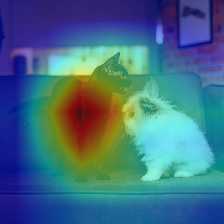The model classifies the image as `Persian cat` by looking at the cat. For this tutorial, we will refer to visualizations like this example as saliency maps, which we define to be heatmaps that highlight pixels influencing the final prediction. There are two types of saliency maps:

1. Model-agnostic Saliency Maps (often called “black-box” methods): These approaches do not need access to the model weights. In general, these methods change the image and observe the changed image’s impact on accuracy. For example, you might remove the center of the image (pictured following). The intuition is: If the image classifier now misclassifies the image, the image center must have been important. We can repeat this and randomly remove parts of the image each time. In this way, we can produce a heatmap like previously, by highlighting the patches that damaged accuracy the most.1. Model-aware Saliency Maps (often called “white-box” methods): These approaches require access to the model’s weights. We will discuss one such method in more detail in the next section.

This concludes our brief overview of saliency maps. In the next step, you will implement one model-aware technique called a Class Activation Map (CAM).

## Step 3 — Generating Class Activation Maps (CAM)

Class Activation Maps (CAMs) are a type of model-aware saliency method. To understand how a CAM is computed, we first need to discuss what the last few layers in a classification network do. Following is an illustration of a typical image-classification neural network, for the method in this paper on Learning Deep Features for Discriminative Localization.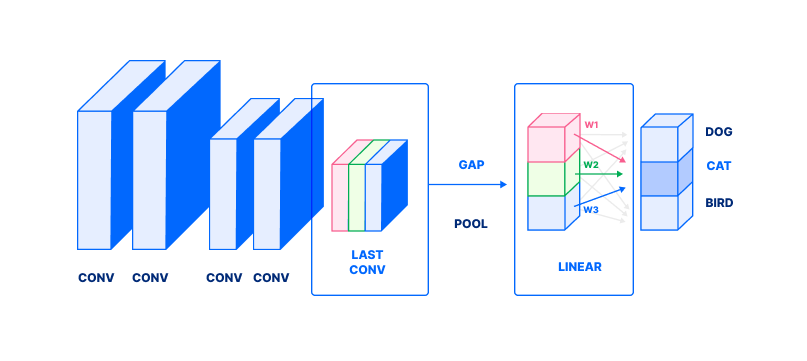The figure describes the following process in a classification neural network. Note the image is represented as a stack of rectangles; for a refresher on how images are represented as a tensor, see How to Build an Emotion-Based Dog Filter in Python 3 (Step 4):

1. Focus on the second-to-last layer’s outputs, labeled LAST CONV with blue, red, and green rectangles.
2. This output undergoes a global average pool (denoted as GAP). GAP averages values in each channel (colored rectangle) to produce a single value (corresponding colored box, in LINEAR).
3. Finally, those values are combined in a weighted sum (with weights denoted by w1, w2, w3) to produce a probability (dark gray box) of a class. In this case, these weights correspond to CAT. In essence, each wi answers: “How important is the ith channel to detecting a Cat?”
4. Repeat for all classes (light gray circles) to obtain probabilities for all classes.

We’ve omitted several details that are not necessary to explain CAM. Now, we can use this to compute CAM. Let us revisit an expanded version of this figure, still for the method in the same paper. Focus on the second row.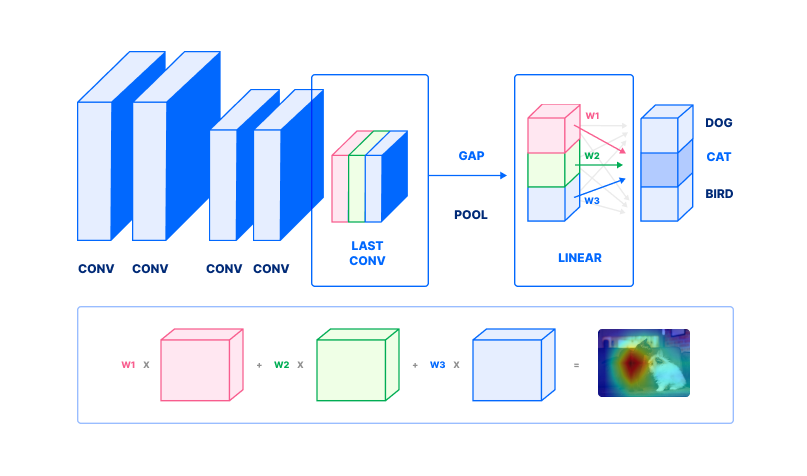1. To compute a class activation map, take the second-to-last layer’s outputs. This is depicted in the second row, outlined by blue, red, and green rectangles corresponding to the same colored rectangles in the first row.
2. Pick a class. In this case, we pick “Australian Terrier”. Find the weights w1, w2wn corresponding to that class.
3. Each channel (colored rectangle) is then weighted by w1, w2wn. Note we do not perform a global average pool (step 2 from the previous figure). Compute the weighted sum, to obtain a class activation map (far right, second row in figure).

This final weighted sum is the class activation map.

Next, we will implement class activation maps. This section will be broken into the three steps that we’ve already discussed:

1. Take the second-to-last layer’s outputs.
2. Find weights `w1`, `w2``wn`.
3. Compute a weighted sum of outputs.

Start by creating a new file `step_3_cam.py`:

``````nano step_3_cam.py

``````

First, add the Python boilerplate; import the necessary packages and declare a `main` function:

step_3_cam.py
``````"""Generate Class Activation Maps"""
import numpy as np
import sys
import torch
import torchvision.models as models
import torchvision.transforms as transforms
import matplotlib.cm as cm

from PIL import Image

def main():
pass

if __name__ == '__main__':
main()
``````

Create an image loader that will load, resize, and crop your image, but leave the color untouched. This ensures your image has the correct dimensions. Add this before your `main` function:

step_3_cam.py
``````. . .
"""Load raw 224x224 center crop of image"""
image = Image.open(sys.argv)
transform = transforms.Compose([
transforms.Resize(224),  # resize smaller side of image to 224
transforms.CenterCrop(224),  # take center 224x224 crop
])
return transform(image)
. . .
``````

In `load_raw_image`, you first access the one argument passed to the script `sys.argv`. Then, open the image specified using `Image.open`. Next, you define a number of different transformations to apply to the images that are passed to your neural network:

• `transforms.Resize(224)`: Resizes the smaller side of the image to 224. For example, if your image is 448 x 672, this operation would downsample the image to 224 x 336.
• `transforms.CenterCrop(224)`: Takes a crop from the center of the image, of size 224 x 224.
• `transform(image)`: Applies the sequence of image transformations defined in the previous lines.

Next, load the pretrained model. Add this function after your first `load_raw_image` function, but before the `main` function:

step_3_cam.py
``````. . .
def get_model():
"""Get model, set forward hook to save second-to-last layer's output"""
net = models.resnet18(pretrained=True).eval()
layer = net.layer4.conv2

def store_feature_map(self, _, output):
self._parameters['out'] = output
layer.register_forward_hook(store_feature_map)

return net, layer
. . .
``````

In the `get_model` function, you:

1. Instantiate a pretrained model `models.resnet18(pretrained=True)`.
2. Change the model’s inference mode to eval by calling `.eval()`.
3. Define `layer...`, the second-to-last layer, which we will use later.
4. Add a “forward hook” function. This function will save the layer’s output when the layer is executed. We do this in two steps, first defining a `store_feature_map` hook and then binding the hook with `register_forward_hook`.
5. Return both the network and the second-to-last layer.

Next, compute the class activation map itself. Add this function before your `main` function:

step_3_cam.py
``````. . .
def compute_cam(net, layer, pred):
"""Compute class activation maps

:param net: network that ran inference
:param layer: layer to compute cam on
:param int pred: prediction to compute cam for
"""

# 1. get second-to-last-layer output
features = layer._parameters['out']

# 2. get weights w_1, w_2, ... w_n
weights = net.fc._parameters['weight'][pred]

# 3. compute weighted sum of output
cam = (features.T * weights).sum(2)

# normalize cam
cam -= cam.min()
cam /= cam.max()
cam = cam.detach().numpy()
return cam
. . .
``````

The `compute_cam` function mirrors the three steps outlined at the start of this section and in the section before.

1. Take the second-to-last layer’s outputs, using the feature maps our forward hook saved in `layer._parameters`.
2. Find weights `w1`, `w2``wn` in the final linear layer `net.fc_parameters['weight']`. Access the `pred`th row of weights, to obtain weights for our predicted class.
3. Compute a weighted sum of outputs. `(features.T * weights).sum(...)`. The argument `2` means we compute a sum along the index `2` dimension of the provided tensor.
4. Normalize the class activation map, so that all values fall in between 0 and 1—`cam -= cam.min(); cam /= cam.max()`.
5. Detach the PyTorch tensor from the computation graph `.detach()`. Convert the CAM from a PyTorch tensor object into a numpy array. `.numpy()`.

This concludes computation for a class activation map.

Our last helper function is a utility that saves the class activation map. Add this function before your `main` function:

step_3_cam.py
``````. . .
def save_cam(cam):
# save heatmap
heatmap = (cm.jet_r(cam) * 255.0)[..., 2::-1].astype(np.uint8)
heatmap = Image.fromarray(heatmap).resize((224, 224))
heatmap.save('heatmap.jpg')
print(' * Wrote heatmap to heatmap.jpg')

# save heatmap on image
combined = (np.array(image) * 0.5 + np.array(heatmap) * 0.5).astype(np.uint8)
Image.fromarray(combined).save('combined.jpg')
print(' * Wrote heatmap on image to combined.jpg')
. . .
``````

This utility `save_cam` performs the following:

1. Colorize the heatmap `cm.jet_r(cam)`. The output is in the range `[0, 1]` so multiply by `255.0`. Furthermore, the output (1) contains a 4th alpha channel and (2) the color channels are ordered as BGR. We use indexing `[..., 2::-1]` to solve both problems, dropping the alpha channel and inverting the color channel order to be RGB. Finally, cast to unsigned integers.
2. Convert the image `Image.fromarray` into a PIL image and use the image’s image-resize utility `.resize(...)`, then the `.save(...)` utility.
3. Load a raw image, using the utility `load_raw_image` we wrote earlier.
4. Superimpose the heatmap on top of the image by adding `0.5` weight of each. Like before, cast the result to unsigned integers `.astype(...)`.
5. Finally, convert the image into PIL, and save.

Next, populate the main function with some code to run the neural network on a provided image:

step_3_cam.py
``````. . .
def main():
"""Generate CAM for network's predicted class"""
net, layer = get_model()

out = net(x)
_, (pred,) = torch.max(out, 1)  # get class with highest probability

cam = compute_cam(net, layer, pred)
save_cam(cam)
. . .
``````

In `main`, run the network to obtain a prediction.

2. Fetch the pretrained neural network.
3. Run the neural network on the image.
4. Find the highest probability with `torch.max`. `pred` is now a number with the index of the most likely class.
5. Compute the CAM using `compute_cam`.
6. Finally, save the CAM using `save_cam`.

This now concludes our class activation script. Save and close your file. Check that your script matches the `step_3_cam.py` in this repository.

Then, run the script:

``````python step_3_cam.py assets/catdog.jpg

``````

Your script will output the following:

``````Output * Wrote heatmap to heatmap.jpg
* Wrote heatmap on image to combined.jpg
``````

This will produce a `heatmap.jpg` and `combined.jpg` akin to the following images showing the heatmap and the heatmap combined with the cat/dog image.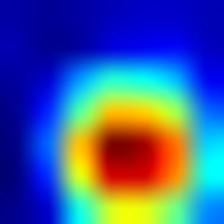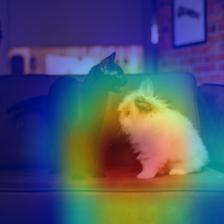You have produced your first saliency map. We will end the article with more links and resources for generating other kinds of saliency maps. In the meantime, let us now explore a second approach to explainability—namely, making the model itself interpretable.

## Step 4 — Using Neural-Backed Decision Trees

Decision Trees belong to a family of rule-based models. A decision tree is a data tree that displays possible decision pathways. Each prediction is the result of a series of predictions.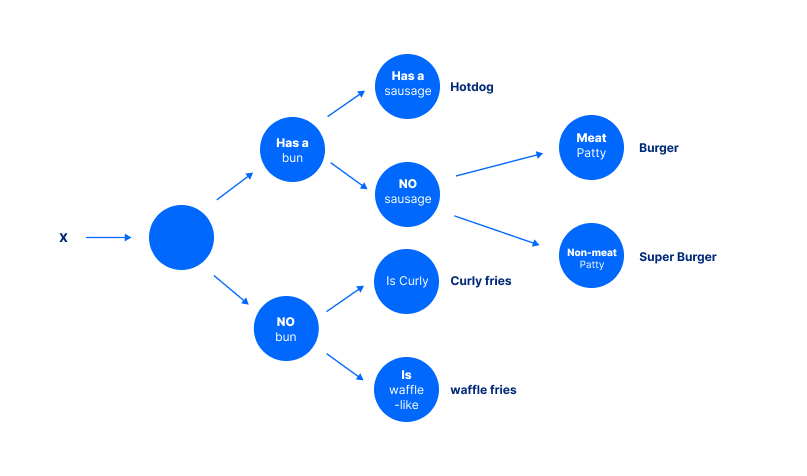Instead of just outputting a prediction, each prediction also comes with justification. For example, to arrive at the conclusion of “Hotdog” for this figure the model must first ask: “Does it have a bun?”, then ask: “Does it have a sausage?” Each of these intermediate decisions can be verified or challenged separately. As a result, classic machine learning calls these rule-based systems “interpretable.”

One question is: How are these rules created? Decision Trees warrant a far more detailed discussion of its own but in short, rules are created to “split classes as much as possible.” Formally, this is “maximizing information gain.” In the limit, maximizing this split makes sense: If the rules perfectly split classes, then our final predictions will always be correct.

Now, we move on to using a neural network and decision tree hybrid. For more on decision trees, see Classification and Regression Trees (CART) overview.

Now, we will run inference on a neural network and decision tree hybrid. As we will find, this gives us a different type of explainability: direct-model interpretability.

Start by creating a new file called `step_4_nbdt.py`:

``````nano step_4_nbdt.py

``````

First, add the Python boilerplate. Import the necessary packages and declare a `main` function. `maybe_install_wordnet` sets up a prerequisite that our program may need:

step_4_nbdt.py
``````"""Run evaluation on a single image, using an NBDT"""

from nbdt.model import SoftNBDT, HardNBDT
from pytorchcv.models.wrn_cifar import wrn28_10_cifar10
from torchvision import transforms
from nbdt.utils import DATASET_TO_CLASSES, load_image_from_path, maybe_install_wordnet
import sys

maybe_install_wordnet()

def main():
pass

if __name__ == '__main__':
main()
``````

Start by loading the pretrained model, as before. Add the following before your `main` function:

step_4_nbdt.py
``````. . .
def get_model():
model = wrn28_10_cifar10()
model = HardNBDT(
pretrained=True,
dataset='CIFAR10',
arch='wrn28_10_cifar10',
model=model)
return model
. . .
``````

This function does the following:

1. Creates a new model called WideResNet `wrn28_10_cifar10()`.
2. Next, it creates the neural-backed decision tree variant of that model, by wrapping it with `HardNBDT(..., model=model)`.

Next, load and preprocess the image for model inference. Add the following before your `main` function:

step_4_nbdt.py
``````. . .
assert len(sys.argv) > 1, "Need to pass image URL or image path as argument"
transform = transforms.Compose([
transforms.Resize(32),
transforms.CenterCrop(32),
transforms.ToTensor(),
transforms.Normalize((0.4914, 0.4822, 0.4465), (0.2023, 0.1994, 0.2010)),
])
x = transform(im)[None]
return x
. . .
``````

In `load_image`, you start by loading the image from the provided URL, using a custom utility method called `load_image_from_path`. Next, you define a number of different transformations to apply to the images that are passed to your neural network:

• `transforms.Resize(32)`: Resizes the smaller side of the image to 32. For example, if your image is 448 x 672, this operation would downsample the image to 32 x 48.
• `transforms.CenterCrop(224)`: Takes a crop from the center of the image, of size 32 x 32.
• `transforms.ToTensor()`: Converts the image into a PyTorch tensor. All PyTorch models require PyTorch tensors as input.
• `transforms.Normalize(mean=..., std=...)`: Standardizes your input by subtracting the mean, then dividing by the standard deviation. This is described more precisely in the torchvision documentation.

Finally, apply the image transformations to the image `transform(im)[None]`.

Next, define a utility function to log both the prediction and the intermediate decisions that led up to it. Place this before your `main` function:

step_4_nbdt.py
``````. . .
def print_explanation(outputs, decisions):
"""Print the prediction and decisions"""
_, predicted = outputs.max(1)
cls = DATASET_TO_CLASSES['CIFAR10'][predicted]
print('Prediction:', cls, '// Decisions:', ', '.join([
'{} ({:.2f}%)'.format(info['name'], info['prob'] * 100) for info in decisions
][1:]))  # [1:] to skip the root
. . .
``````

The `print_explanations` function computes and logs predictions and decisions:

1. Starts by computing the index of the highest probability class `outputs.max(1)`.
2. Then, it converts that prediction into a human readable class name using the dictionary `DATASET_TO_CLASSES['CIFAR10'][predicted]`.
3. Finally, it prints the prediction `cls` and the decisions `info['name'], info['prob']...`.

Conclude the script by populating the `main` with utilities we have written so far:

step_4_nbdt.py
``````. . .
def main():
model = get_model()
outputs, decisions = model.forward_with_decisions(x)  # use `model(x)` to obtain just logits
print_explanation(outputs, decisions)
``````

We perform model inference with explanations in several steps:

1. Load the model `get_model`.
2. Load the image `load_image`.
3. Run model inference `model.forward_with_decisions`.
4. Finally, print the prediction and explanations `print_explanations`.

Close your file, and double-check your file contents matches `step_4_nbdt.py`. Then, run your script on the photo from earlier of two pets side-by-side.

``````python step_4_nbdt.py assets/catdog.jpg

``````

This will output the following, both the prediction and the corresponding justifications.

``````OutputPrediction: cat // Decisions: animal (99.34%), chordate (92.79%), carnivore (99.15%), cat (99.53%)
``````

This concludes the neural-backed decision tree section.

## Conclusion

You have now run two types of Explainable AI approaches: a post-hoc explanation like saliency maps and a modified interpretable model using a rule-based system.

There are many explainability techniques not covered in this tutorial. For further reading, please be sure to check out other ways to visualize and interpret neural networks; the utilities number many, from debugging to debiasing to avoiding catastrophic errors. There are many applications for Explainable AI (XAI), from sensitive applications like medicine to other mission-critical systems in self-driving cars.

]]>
Alvin Wan 2020-11-23T19:21:07.559Z
<![CDATA[An Introduction to Computer Vision in JavaScript using OpenCV.js]]> tag:www.digitalocean.com,2005:/community/tutorials/introduction-to-computer-vision-in-javascript-using-opencvjs 2020-10-20T19:26:54.591Z Introduction

OpenCV, or Open Source Computer Vision Library, is a powerful library used for image processing and image recognition. The library has a massive community and has been used extensively in many fields, from face detection to interactive art. It was first built in C++, but bindings have been created for different languages, such as Python and Java. It is even available in JavaScript as OpenCV.js, which is what we’ll be using for this tutorial.

In this project, we will create a webpage where a user can upload an image in order to detect all the circles contained in it. We will highlight the circles with a black outline, and the user will be able to download the modified image.

The code for this project is available in this GitHub repo.

## Prerequisites

To complete this tutorial, you will need to pull in the OpenCV.js library. The 3.3.1 version is available here:

``````https://docs.opencv.org/3.3.1/opencv.js
``````

Save this file locally as `opencv.js` in a place where you can easily locate it.

## Step 1 — Setting Up the Project

To get started, you will first need to create a space for your project. Create a directory named `opencvjs-project`:

``````mkdir opencvjs-project
``````

Move your local copy of `opencv.js` to this directory.

Next, add an `index.html` file with the following template:

index.html
``````<!DOCTYPE html>
<html>
<title>OpenCV.js</title>
<body>

<!-- Our HTML will go here-->

<script type="text/javascript">
// Our JavaScript code will go here
</script>

</body>
</html>
``````

In addition to the existing empty `<script>` tag in this file, add a new `<script>` tag which references the local `opencv.js` file. The script is quite large and takes a bit of time to load, so it is better load it asynchronously. This can be done by adding `async` to the `<script>` tag:

index.html
``````  <script type="text/javascript">
// Our JavaScript code will go here
</script>
<script async src="opencv.js" type="text/javascript"></script>
``````

As OpenCV.js may not be ready immediately due to the file size, we can provide a better user experience by showing that the content is being loaded. We can add a loading spinner to the page (credit to Sampson on StackOverflow).

First, add a `<div>` element `<body>`:

index.html
``````<body>

<!-- Our HTML will go here-->
<div class="modal"></div>

<script type="text/javascript">
// Our JavaScript code will go here
</script>
<script async src="opencv.js" type="text/javascript"></script>

</body>
``````

Next, add the following CSS into a separate `<style>` tag in the `<head>` of `index.html`. The spinner is invisible by default (thanks to `display: none;`):

index.html
``````/* display loading gif and hide webpage */
.modal {
display:    none;
position:   fixed;
z-index:    1000;
top:        0;
left:       0;
height:     100%;
width:      100%;
background: rgba( 255, 255, 255, .8)
url('http://i.stack.imgur.com/FhHRx.gif')
50% 50%
no-repeat;
}

/* prevent scrollbar from display during load */
overflow: hidden;
}

display: block;
}
``````

To show the loading gif, we can add the `"loading"` class to the body. Add the following to the empty `<script>`.

index.html
``````document.body.classList.add('loading');
``````

When OpenCV.js loads, we’ll want to hide the loading gif. Modify the `<script>` tag which references the local `opencv.js` file to add an `onload` event listener:

index.html
``````<script async src="opencv.js" onload="onOpenCvReady();" type="text/javascript"></script>
``````

Then add `onOpenCvReady` to the other `<script>` tag to handle removing the `"loading"` class:

index.html
``````// previous code is here

}
``````

Open the HTML page in your browser and check that OpenCV.js loads as expected.

Note: Using your browser’s developer tools, you should verify that there are no error messages in the Console tab and that the Network tab shows the `opencv.js` file being referenced properly. You will be periodically refreshing this page in your browser to view your latest changes.

Now that you’ve set up the project, you’re ready to build the image upload functionality.

To create the upload functionality, start by adding in an `<input>` element to `index.html`:

index.html
``````<input type="file" id="fileInput" name="file" />
``````

If we just want to display the source image, we’ll also need to add an `<img>` element and an event listener, which responds to change on the `<input>` element. Copy the following tag and place it under the `<input>` tag:

index.html
``````<img id="imageSrc" alt="No Image" />
``````

Get both the `<img>` element and the `<input>` element using their `id` values:

index.html
``````// previous code is here

let imgElement = document.getElementById('imageSrc');
let inputElement = document.getElementById('fileInput');
``````

Now, add the event listener, which triggers when the `<input>` changes (i.e., when a file is uploaded). From the change event, it’s possible to access the uploaded file (`event.target.files`), and convert it into a URL using `URL.createObjectURL`). The image’s `src` attribute can be updated to this URL:

index.html
``````// previous code is here

inputElement.onchange = function() {
imgElement.src = URL.createObjectURL(event.target.files);
};
``````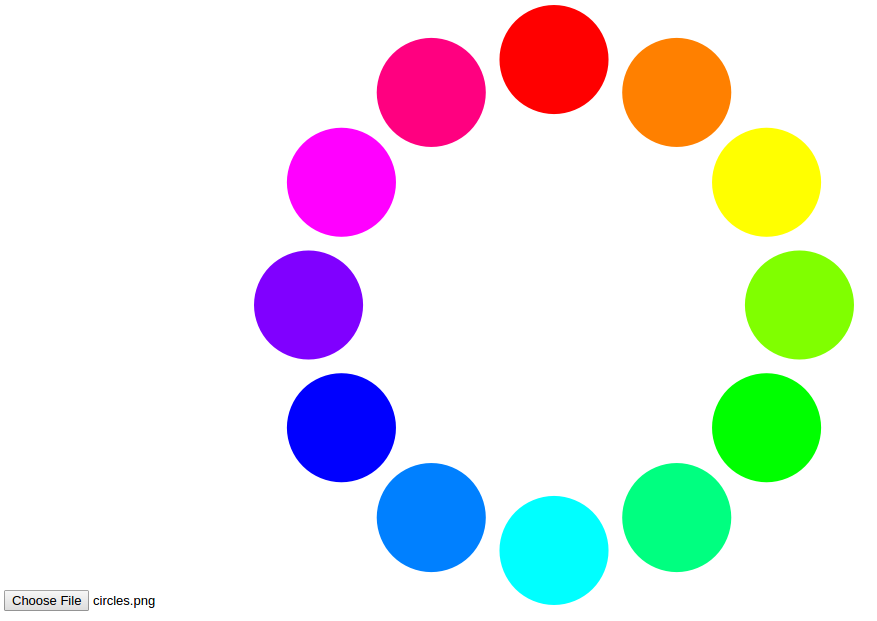Next to the original image, we can display a second image indicating the detected circles. The image will be displayed with a `<canvas>` element, which is used for drawing graphics with JavaScript:

index.html
``````<canvas id="imageCanvas"></canvas>
``````

We can add another event listener which updates the `<canvas>` with the uploaded image:

index.html
``````// previous code is here

cv.imshow('imageCanvas', image);
image.delete();
};
``````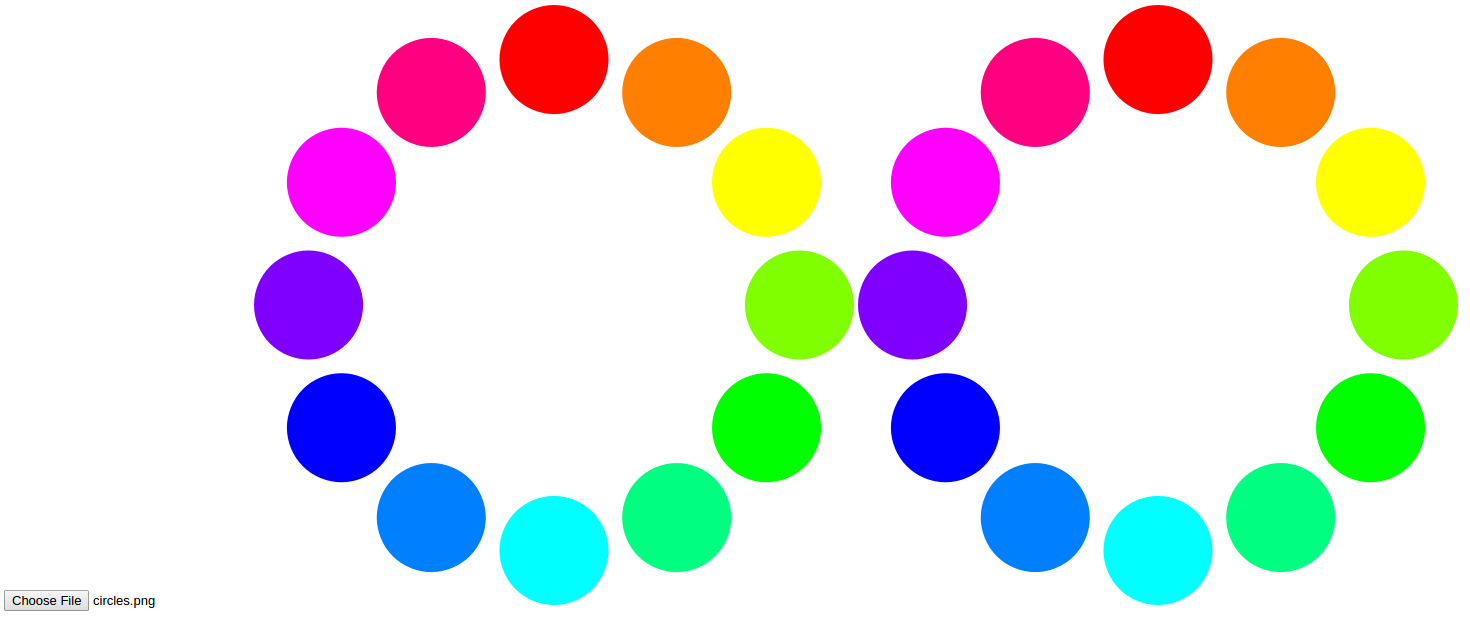In this step, you’ve set up the image upload and display functionality. In the next step, you’ll explore how to use OpenCV to detect circles.

## Step 3 — Detecting Circles

This is where the power of OpenCV is evident, as detecting circles is a built-in task. We want to find the circles when the user clicks a button, so we’ll need to add the button and an event listener:

index.html
``````<button type="button" id="circlesButton" class="btn btn-primary">Circle Detection</button>
``````
index.html
``````// previous code is here

document.getElementById('circlesButton').onclick = function() {
// circle detection code
};
``````

Depending on the image, circle detection may take a while, so it is a good idea to disable the button to prevent the user from hitting it multiple times. It could also be useful to show a loading spinner on the button. We can reuse the loading gif from the initial script load:

index.html
``````// previous code is here

document.getElementById('circlesButton').onclick = function() {
this.disabled = true;

// circle detection code

this.disabled = false;
};
``````

The first step to detecting the circles is reading the image from the `<canvas>`.

In OpenCV, images are stored and manipulated as `Mat` objects. These are essentially matrices that hold values for each pixel in the image.

For our circle detection, we’re going to need three Mat objects:

• `srcMat` - Holds the source image (from which circles are detected)
• `circlesMat` - Stores the circles we detect
• `displayMatOne` - Displays to the user (on which we will draw our highlighted circles)

For the final `Mat`, we can make a copy of the first using the `clone` function:

index.html
``````// circle detection code
let displayMat = srcMat.clone();
let circlesMat = new cv.Mat();
``````

The `srcMat` needs to be converted to grayscale. This makes circle detection faster by simplifying the image. We can use `cvtColor` function to do this.

This function needs:

• the source `Mat` (`srcMat`)
• the destination `Mat` (in this case, the source and the destination Mat will be the same `srcMat`)
• a value which refers to the color conversion. `cv.COLOR_RGBA2GRAY` is the constant for grayscale.
index.html
``````cv.cvtColor(srcMat, srcMat, cv.COLOR_RGBA2GRAY);
``````

The `cvtColor` function, like other OpenCV.js functions, accepts more parameters. These are not required, so they will be set to the default. You can refer to the documentation for better customization.

Once the image is converted to grayscale, it’s possible to use the `HoughCircles` function to detect the circles.

This function needs:

• a source `Mat` from where it’ll find the circles (`srcMat`)
• a destination `Mat` where it’ll store the circles (`circlesMat`)
• the method to detect circles (`cv.HOUGH_GRADIENT`)
• the inverse ratio of the accumulator resolution (`1`)
• the minimum distance between the center point of circles (`45`)

There are more parameters, thresholds for the algorithm (`75` and `40`), which can be played with to improve accuracy for your images.

It is also possible to limit the range of the circles you want to detect by setting a minimum (`0`) and maximum radius (`0`).

index.html
``````cv.HoughCircles(srcMat, circlesMat, cv.HOUGH_GRADIENT, 1, 45, 75, 40, 0, 0);
``````

Now, we should have a `Mat` object with the circles detected in it.

Next, we’ll draw the circles in our `<canvas>`.

## Step 4 — Drawing Circles

All the circles which were detected can now be highlighted. We want to make an outline around each circle to show to the user. To draw a circle with OpenCV.js, we need the center point and the radius. These values are stored inside `circlesMat`, so we can retrieve it by looping through the matrix’s columns:

index.html
``````for (let i = 0; i < circlesMat.cols; ++i) {
// draw circles
}
``````

The `circlesMat` stores the `x` and `y` values for the center point and the radius sequentially.

For example, for the first circle, it would be possible to retrieve the values as follows:

``````let x = circlesMat.data32F;
let y = circlesMat.data32F;
``````

To get all the values for each circle, we can do the following:

index.html
``````for (let i = 0; i < circlesMat.cols; ++i) {
let x = circlesMat.data32F[i * 3];
let y = circlesMat.data32F[i * 3 + 1];
let radius = circlesMat.data32F[i * 3 + 2];

// draw circles
}
``````

Finally, with all these values, we are able to draw outlines around the circles.

To draw circles in OpenCV.js, we need:

• a destination `Mat` (the image we’re going to display to the user - `displayMat`)
• a center `Point` (using the x and y values)
• a scalar (an array of RGB values)

There are also additional parameters which can be passed into `circles`, such as the line thickness, which for in this example is `3`:

index.html
``````let center = new cv.Point(x, y);
cv.circle(displayMat, center, radius, [0, 0, 0, 255], 3);
``````

All the code for drawing circles is as follows:

index.html
``````for (let i = 0; i < circlesMat.cols; ++i) {
let x = circlesMat.data32F[i * 3];
let y = circlesMat.data32F[i * 3 + 1];
let radius = circlesMat.data32F[i * 3 + 2];
let center = new cv.Point(x, y);

// draw circles
cv.circle(displayMat, center, radius, [0, 0, 0, 255], 3);
}
``````

Once we’re done drawing all the circles on `displayMat`, we can display it to the user:

index.html
``````cv.imshow('imageCanvas', displayMat);
``````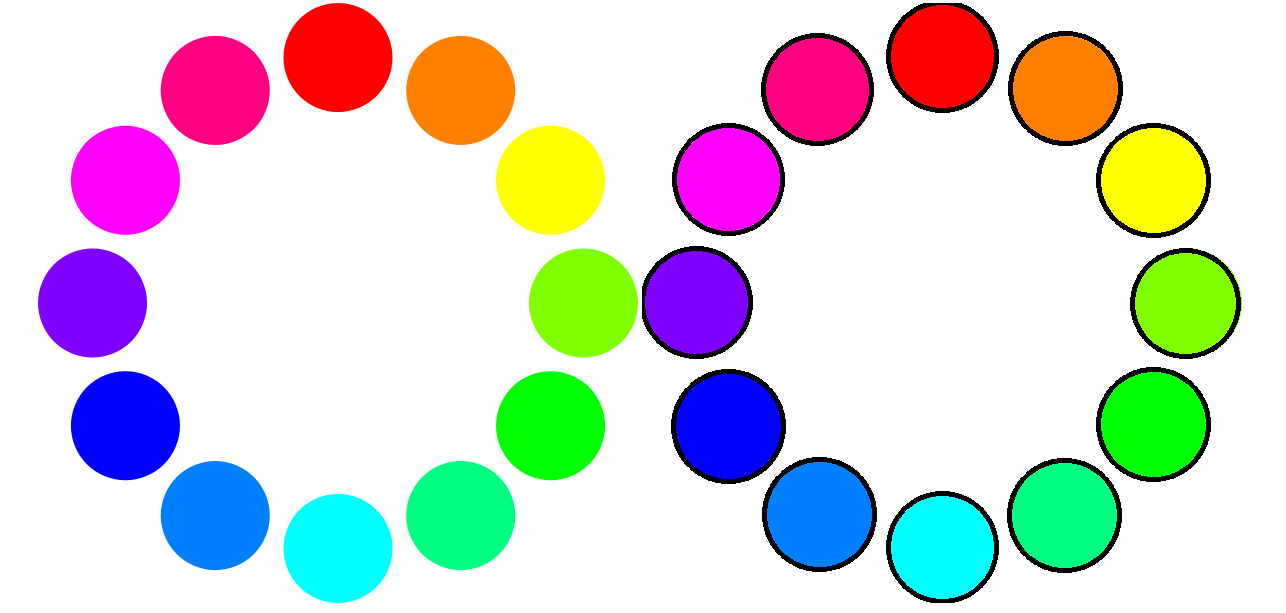Finally, it’s good practice to clean up the `Mat` objects which we’ll no longer be needing. This is done to prevent memory problems:

index.html
``````srcMat.delete();
displayMat.delete();
circlesMat.delete();
``````

When we put it all together, the circle detection and drawing code will look like this:

index.html
``````// previous code is here

document.getElementById('circlesButton').onclick = function() {
this.disabled = true;

let displayMat = srcMat.clone();
let circlesMat = new cv.Mat();

cv.cvtColor(srcMat, srcMat, cv.COLOR_RGBA2GRAY);

cv.HoughCircles(srcMat, circlesMat, cv.HOUGH_GRADIENT, 1, 45, 75, 40, 0, 0);

for (let i = 0; i < circlesMat.cols; ++i) {
let x = circlesMat.data32F[i * 3];
let y = circlesMat.data32F[i * 3 + 1];
let radius = circlesMat.data32F[i * 3 + 2];
let center = new cv.Point(x, y);

// draw circles
cv.circle(displayMat, center, radius, [0, 0, 0, 255], 3);
}

cv.imshow('imageCanvas', displayMat);

srcMat.delete();
displayMat.delete();
circlesMat.delete();

this.disabled = false;
};
``````

With that, you have added the logic to detect and draw circles around circles in images.

After the image has been modified, the user may want to download it. To do this, add a hyperlink to your `index.html` file:

index.html
``````<a href="#" id="downloadButton">Download Image</a>
``````

We set the `href` to the image URL and the `download` attribute to the image filename. Setting the `download` attribute indicates to the browser that the resource should be downloaded rather than navigating to it. We can create the image URL from the `<canvas>` using the function `toDataURL()`.

Add the following JavaScript to the bottom of the `<script>`:

index.html
``````// previous code is here

this.href = document.getElementById('imageCanvas').toDataURL();
};
``````

## Conclusion

Detecting circles is possible with OpenCV. Once you get accustomed to manipulating images as `Mat` objects, there is so much more you can do. The `HoughCircles` algorithm is one of many provided by OpenCV to make image processing and image recognition that much easier.

You can find more tutorials, including face recognition and template matching, on the OpenCV website. You can also read more about computer vision by visiting the machine learning topic page.

]]>
Lily Rae 2020-10-20T19:26:54.591Z
<![CDATA[How do I load locally stored tweets instead of using from nltk.corpus import twitter_samples.]]> tag:www.digitalocean.com,2005:/community/questions/how-do-i-load-locally-stored-tweets-instead-of-using-from-nltk-corpus-import-twitter_samples 2020-08-06T16:26:51.090Z Every example I have seen on the internet about sentiment analysis uses a pre-existing sample file imported from nltk.corpus but I already have multiple .db files for training. How would I use those and not import from nltk.corpus? Anyone?

]]>
ctoshpokh 2020-08-06T16:26:51.090Z
<![CDATA[How To Trick a Neural Network in Python 3]]> tag:www.digitalocean.com,2005:/community/tutorials/how-to-trick-a-neural-network-in-python-3 2020-07-30T19:30:37.088Z The author selected Dev Color to receive a donation as part of the Write for DOnations program.

Could a neural network for animal classification be fooled? Fooling an animal classifier may have few consequences, but what if our face authenticator could be fooled? Or our self-driving car prototype’s software? Fortunately, legions of engineers and research stand between a prototype computer-vision model and production-quality models on our mobile devices or cars. Still, these risks have significant implications and are important to consider as a machine-learning practitioner.

In this tutorial, you will try “fooling” or tricking an animal classifier. As you work through the tutorial, you’ll use `OpenCV`, a computer-vision library, and `PyTorch`, a deep learning library. You will cover the following topics in the associated field of adversarial machine learning:

• Create a targeted adversarial example. Pick an image, say, of a dog. Pick a target class, say, a cat. Your goal is to trick the neural network into believing the pictured dog is a cat.
• Create an adversarial defense. In short, protect your neural network against these tricky images, without knowing what the trick is.

By the end of the tutorial, you will have a tool for tricking neural networks and an understanding of how to defend against tricks.

## Prerequisites

To complete this tutorial, you will need the following:

## Step 1 — Creating Your Project and Installing Dependencies

Let’s create a workspace for this project and install the dependencies you’ll need. You’ll call your workspace `AdversarialML`:

``````mkdir ~/AdversarialML

``````

Navigate to the `AdversarialML` directory:

``````cd ~/AdversarialML

``````

Make a directory to hold all your assets:

``````mkdir ~/AdversarialML/assets

``````

Then create a new virtual environment for the project:

``````python3 -m venv adversarialml

``````

``````source adversarialml/bin/activate

``````

Then install PyTorch, a deep-learning framework for Python that you’ll use in this tutorial.

On macOS, install Pytorch with the following command:

``````python -m pip install torch==1.2.0 torchvision==0.4.0

``````

On Linux and Windows, use the following commands for a CPU-only build:

``````pip install torch==1.2.0+cpu torchvision==0.4.0+cpu -f https://download.pytorch.org/whl/torch_stable.html
pip install torchvision

``````

Now install prepackaged binaries for `OpenCV` and `numpy`, which are libraries for computer vision and linear algebra, respectively. `OpenCV` offers utilities such as image rotations, and `numpy` offers linear algebra utilities such as a matrix inversion:

``````python -m pip install opencv-python==3.4.3.18 numpy==1.14.5

``````

On Linux distributions, you will need to install `libSM.so`:

``````sudo apt-get install libsm6 libxext6 libxrender-dev

``````

With the dependencies installed, let’s run an animal classifier called ResNet18, which we describe next.

## Step 2 — Running a Pretrained Animal Classifier

The `torchvision` library, the official computer vision library for PyTorch, contains pretrained versions of commonly used computer vision neural networks. These neural networks are all trained on ImageNet 2012, a dataset of 1.2 million training images with 1000 classes. These classes include vehicles, places, and most importantly, animals. In this step, you will run one of these pretrained neural networks, called ResNet18. We will refer to ResNet18 trained on ImageNet as an “animal classifier”.

What is ResNet18? ResNet18 is the smallest neural network in a family of neural networks called residual neural networks, developed by MSR (He et al.). In short, He found that a neural network (denoted as a function `f`, with input `x`, and output `f(x)`) would perform better with a “residual connection” `x + f(x)`. This residual connection is used prolifically in state-of-the-art neural networks, even today. For example, FBNetV2, FBNetV3.

``````wget -O assets/dog.jpg https://assets.digitalocean.com/articles/trick_neural_network/step2a.png

````````````wget -O assets/imagenet_idx_to_label.json https://raw.githubusercontent.com/do-community/tricking-neural-networks/master/utils/imagenet_idx_to_label.json

``````

Next, create a script to run your pretrained model on the dog image. Create a new file called `step_2_pretrained.py`:

``````nano step_2_pretrained.py

``````

First, add the Python boilerplate by importing the necessary packages and declaring a `main` function:

step_2_pretrained.py
``````from PIL import Image
import json
import torchvision.models as models
import torchvision.transforms as transforms
import torch
import sys

def main():
pass

if __name__ == '__main__':
main()
``````

Next, load the mapping from neural network output to human-readable class names. Add this directly after your import statements and before your `main` function:

step_2_pretrained.py
``````. . .
def get_idx_to_label():
with open("assets/imagenet_idx_to_label.json") as f:
. . .
``````

Create an image transformation function that will ensure your input image firstly has the correct dimensions, and secondly is normalized correctly. Add the following function directly after the last:

step_2_pretrained.py
``````. . .
def get_image_transform():
transform = transforms.Compose([
transforms.Resize(224),
transforms.CenterCrop(224),
transforms.ToTensor(),
transforms.Normalize(mean=[0.485, 0.456, 0.406],
std=[0.229, 0.224, 0.225])
])
return transform
. . .
``````

In `get_image_transform`, you define a number of different transformations to apply to the images that are passed to your neural network:

• `transforms.Resize(224)`: Resizes the smaller side of the image to 224. For example, if your image is 448 x 672, this operation would downsample the image to 224 x 336.
• `transforms.CenterCrop(224)`: Takes a crop from the center of the image, of size 224 x 224.
• `transforms.ToTensor()`: Converts the image into a PyTorch tensor. All PyTorch models require PyTorch tensors as input.
• `transforms.Normalize(mean=..., std=...)`: Standardizes your input by subtracting the mean, then dividing by the standard deviation. This is described more precisely in the `torchvision` documentation.

Add a utility to predict the animal class, given the image. This method uses both the previous utilities to perform animal classification:

step_2_pretrained.py
``````. . .
def predict(image):
model = models.resnet18(pretrained=True)
model.eval()

out = model(image)

_, pred = torch.max(out, 1)
idx_to_label = get_idx_to_label()
cls = idx_to_label[str(int(pred))]
return cls
. . .
``````

Here the `predict` function classifies the provided image using a pretrained neural network:

• `models.resnet18(pretrained=True)`: Loads a pretrained neural network called ResNet18.
• `model.eval()`: Modifies the model in-place to run in ‘evaluation’ mode. The only other mode is ‘training’ mode, but training mode isn’t needed, as you aren’t training the model (that is, updating the model’s parameters) in this tutorial.
• `out = model(image)`: Runs the neural network on the provided, transformed image.
• `_, pred = torch.max(out, 1)`: The neural network outputs one probability for each possible class. This step computes the index of the class with the highest probability. For example, if `out = [0.4, 0.1, 0.2]`, then `pred = 0`.
• `idx_to_label = get_idx_to_label()`: Obtains a mapping from class index to human-readable class names. For example, the mapping could be `{0: cat, 1: dog, 2: fish}`.
• `cls = idx_to_label[str(int(pred))]`: Convert the predicted class index to a class name. The examples provided in the last two bullet points would yield `cls = idx_to_label = 'cat'`.

step_2_pretrained.py
``````. . .
assert len(sys.argv) > 1, 'Need to pass path to image'
image = Image.open(sys.argv)

transform = get_image_transform()
image = transform(image)[None]
return image
. . .
``````

This will load an image from the path provided in the first argument to the script. `transform(image)[None]` applies the sequence of image transformations defined in the previous lines.

Finally, populate your `main` function with the following, to load your image and classify the animal in the image:

step_2_pretrained.py
``````def main():
print(f'Prediction: {predict(x)}')
``````

Double check that your file matches our final step 2 script at `step_2_pretrained.py` on GitHub. Save and exit your script, and run the animal classifier:

``````python step_2_pretrained.py assets/dog.jpg

``````

This will produce the following output, showing your animal classifier works as expected:

``````OutputPrediction: Pembroke, Pembroke Welsh corgi
``````

That concludes running inference with your pretrained model. Next, you will see an adversarial example in action by tricking a neural network with impercetible differences in the image.

## Step 3 — Trying an Adversarial Example

Now, you will synthesize an adversarial example, and test the neural network on that example. For this tutorial, you will build adversarial examples of the form `x + r`, where `x` is the original image and `r` is some “perturbation”. You will eventually create the perturbation `r` yourself, but in this step, you will download one we created for you beforehand. Start by downloading the perturbation `r`:

``````wget -O assets/adversarial_r.npy https://github.com/do-community/tricking-neural-networks/blob/master/outputs/adversarial_r.npy?raw=true

``````

Now composite the picture with the perturbation. Create a new file called `step_3_adversarial.py`:

``````nano step_3_adversarial.py

``````

In this file, you will perform the following three-step process, to produce an adversarial example:

1. Transform an image
2. Apply the perturbation `r`
3. Inverse transform the perturbed image

At the end of step 3, you will have an adversarial image. First, import the necessary packages and declare a `main` function:

``````from PIL import Image
import torchvision.transforms as transforms
import torch
import numpy as np
import os
import sys

from step_2_pretrained import get_idx_to_label, get_image_transform, predict, load_image

def main():
pass

if __name__ == '__main__':
main()
``````

Next, create an “image transformation” that inverts the earlier image transformation. Place this after your imports, before the `main` function:

``````. . .
def get_inverse_transform():
return transforms.Normalize(
mean=[-0.485/0.229, -0.456/0.224, -0.406/0.255],  # INVERSE normalize images, according to https://pytorch.org/docs/stable/torchvision/models.html
std=[1/0.229, 1/0.224, 1/0.255])
. . .
``````

As before, the `transforms.Normalize` operation subtracts the mean and divides by the standard deviation (that is, for the original image `x`, `y = transforms.Normalize(mean=u, std=o) = (x - u) / o`). You do some algebra and define a new operation that reverses this normalize function (`transforms.Normalize(mean=-u/o, std=1/o) = (y - -u/o) / 1/o = (y + u/o) o = yo + u = x`).

As part of the inverse transformation, add a method that transforms a PyTorch tensor back to a PIL image. Add this following the last function:

``````. . .
def tensor_to_image(tensor):
x = tensor.data.numpy().transpose(1, 2, 0) * 255.
x = np.clip(x, 0, 255)
return Image.fromarray(x.astype(np.uint8))
. . .
``````
• `tensor.data.numpy()` converts the PyTorch tensor into a NumPy array. `.transpose(1, 2, 0)` rearranges `(channels, width, height)` into `(height, width, channels)`. This NumPy array is approximately in the range `(0, 1)`. Finally, multiply by 255 to ensure the image is now in the range `(0, 255)`.
• `np.clip` ensures that all values in the image are between `(0, 255)`.
• `x.astype(np.uint8)` ensures all image values are integers. Finally, `Image.fromarray(...)` creates a PIL image object from the NumPy array.

Then, use these utilities to create the adversarial example with the following:

``````. . .
y = x + r
y = get_inverse_transform()(y)
image = tensor_to_image(y)
return image
. . .
``````

This function generates the adversarial example as described at the start of the section:

1. `y = x + r`. Take your perturbation `r` and add it to the original image `x`.
2. `get_inverse_transform`: Obtain and apply the reverse image transformation you defined several lines earlier.
3. `tensor_to_image`: Finally, convert the PyTorch tensor back to an image object.

Finally, modify your `main` function to load the image, load the adversarial perturbation `r`, apply the perturbation, save the adversarial example to disk, and run prediction on the adversarial example:

``````def main():

# save perturbed image
os.makedirs('outputs', exist_ok=True)

# check prediction is new class
print(f'Old prediction: {predict(x)}')
print(f'New prediction: {predict(x + r)}')
``````

Your completed file should match `step_3_adversarial.py` on GitHub. Save the file, exit the editor, and launch your script with:

``````python step_3_adversarial.py assets/dog.jpg

``````

You’ll see this output:

``````OutputOld prediction: Pembroke, Pembroke Welsh corgi
New prediction: goldfish, Carassius auratus
``````

You’ve now created an adversarial example: tricking the neural network into thinking a corgi is a goldfish. In the next step, you will actually create the perturbation `r` that you used here.

## Step 4 — Understanding an Adversarial Example

For a primer on classification, see “How to Build an Emotion-Based Dog Filter”.

Taking a step back, recall that your classification model outputs a probability for each class. During inference, the model predicts the class with the highest probability. During training, you update the model parameters `t` to maximize the probability of the correct class `y`, given your data `x`.

``````argmax_y P(y|x,t)
``````

However, to generate adversarial examples, you now modify your goal. Instead of finding a class, your goal is now to find a new image, `x`. Take any class other than the correct one. Let us call this new class `w`. Your new objective is to maximize the probability of the wrong class.

``````argmax_x P(w|x)
``````

Note that the neural network weights `t` are missing from the above expression. This is because you now assume the role of the adversary: Someone else has trained and deployed a model. You are only allowed to create adversarial inputs and are not allowed to modify the deployed model. To generate the adversarial example `x`, you can run “training”, except instead of updating the neural network weights, you update the input image with the new objective.

As a reminder, for this tutorial, you assume that the adversarial example is an affine transformation of `x`. In other words, your adversarial example takes the form `x + r` for some `r`. In the next step, you will write a script to generate this `r`.

## Step 5 — Creating an Adversarial Example

In this step, you will learn a perturbation `r`, so that your corgi is misclassified as a goldfish. Create a new file called `step_5_perturb.py`:

``````nano step_5_perturb.py

``````

Import the necessary packages and declare a `main` function:

step_5_perturb.py
``````from torch.autograd import Variable
import torchvision.models as models
import torch.nn as nn
import torch.optim as optim
import numpy as np
import torch
import os

from step_2_pretrained import get_idx_to_label, get_image_transform, predict, load_image

def main():
pass

if __name__ == '__main__':
main()
``````

Directly following your imports and before the `main` function, define two constants:

step_5_perturb.py
``````. . .
TARGET_LABEL = 1
EPSILON = 10 / 255.
. . .
``````

The first constant `TARGET_LABEL` is the class to misclassify the corgi as. In this case, index `1` corresponds to “goldfish”. The second constant `EPSILON` is the maximum amount of perturbation allowed for each image value. This limit is introduced so that the image is imperceptibly altered.

Following your two constants, add a helper function to define a neural network and the perturbation parameter `r`:

step_5_perturb.py
``````. . .
def get_model():
net = models.resnet18(pretrained=True).eval()
r = nn.Parameter(data=torch.zeros(1, 3, 224, 224), requires_grad=True)
return net, r
. . .
``````
• `model.resnet18(pretrained=True)` loads a pretrained neural network called ResNet18, like before. Also like before, you set the model to evaluation mode using `.eval`.
• `nn.Parameter(...)` defines a new perturbation `r`, the size of the input image. The input image is also of size `(1, 3, 224, 224)`. The `requires_grad=True` keyword argument ensures that you can update this perturbation `r` in later lines, in this file.

Next, begin modifying your `main` function. Start by loading the model `net`, loading the inputs `x`, and defining the label `label`:

step_5_perturb.py
``````. . .
def main():
print(f'Target class: {get_idx_to_label()[str(TARGET_LABEL)]}')
net, r = get_model()
labels = Variable(torch.Tensor([TARGET_LABEL])).long()
. . .
``````

Next, define both the criterion and the optimizer in your `main` function. The former tells PyTorch what the objective is—that is, what loss to minimize. The latter tells PyTorch how to train your parameter `r`:

step_5_perturb.py
``````. . .
criterion = nn.CrossEntropyLoss()
optimizer = optim.SGD([r], lr=0.1, momentum=0.1)
. . .
``````

Directly following, add the main training loop for your parameter `r`:

step_5_perturb.py
``````. . .
for i in range(30):
r.data.clamp_(-EPSILON, EPSILON)

outputs = net(x + r)
loss = criterion(outputs, labels)
loss.backward()
optimizer.step()

_, pred = torch.max(outputs, 1)
if i % 5 == 0:
print(f'Loss: {loss.item():.2f} / Class: {get_idx_to_label()[str(int(pred))]}')
. . .
``````

On each iteration of this training loop, you:

• `r.data.clamp_(...)`: Ensure the parameter `r` is small, within `EPSILON` of 0.
• `optimizer.zero_grad()`: Clear any gradients you computed in the previous iteration.
• `model(x + r)`: Run inference on the modified image `x + r`.
• Compute the `loss`.
• Compute the gradient `loss.backward`.
• Take a gradient descent step `optimizer.step`.
• Compute the prediction `pred`.
• Finally, report the loss and predicted class `print(...)`.

Next, save the final perturbation `r`:

step_5_perturb.py
``````def main():
. . .
for i in range(30):
. . .
. . .
``````

Directly following, still in the `main` function, save the perturbed image:

step_5_perturb.py
``````. . .
os.makedirs('outputs', exist_ok=True)
``````

Finally, run prediction on both the original image and the adversarial example:

step_5_perturb.py
``````    print(f'Old prediction: {predict(x)}')
print(f'New prediction: {predict(x + r)}')
``````

Double check your script matches `step_5_perturb.py` on GitHub. Save, exit, and run the script:

``````python step_5_perturb.py assets/dog.jpg

``````

Your script will output the following.

``````OutputTarget class: goldfish, Carassius auratus
Loss: 17.03 / Class: Pembroke, Pembroke Welsh corgi
Loss: 8.19 / Class: Pembroke, Pembroke Welsh corgi
Loss: 5.56 / Class: Pembroke, Pembroke Welsh corgi
Loss: 3.53 / Class: Pembroke, Pembroke Welsh corgi
Loss: 1.99 / Class: Pembroke, Pembroke Welsh corgi
Loss: 1.00 / Class: goldfish, Carassius auratus
Old prediction: Pembroke, Pembroke Welsh corgi
New prediction: goldfish, Carassius auratus
``````

The last two lines indicate you have now completed construction of an adversarial example from scratch. Your neural network now classifies a perfectly reasonable corgi image as a goldfish.

You’ve now shown that neural networks can be fooled easily—what’s more, the lack of robustness to adversarial examples has significant consequences. A natural next question is this: How can you combat adversarial examples? A good amount of research has been conducted by various organizations, including OpenAI. In the next section, you’ll run a defense to thwart this adversarial example.

## Step 6 — Defending Against Adversarial Examples

In this step, you will implement a defense against adversarial examples. The idea is the following: You are now the owner of the animal classifier being deployed to production. You don’t know what adversarial examples may be generated, but you can modify the image or the model to protect against attacks.

Before you defend, you should see for yourself how imperceptible the image manipulation is. Open both of the following images:

1. `assets/dog.jpg`
2. `outputs/adversarial.png`

Here, you show both side by side. Your original image will have a different aspect ratio. Can you tell which is the adversarial example?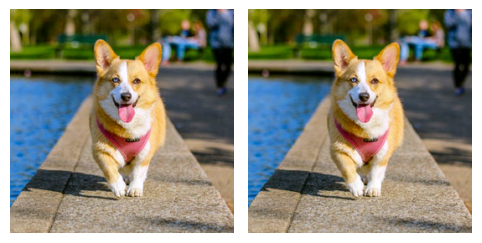Notice that the new image looks identical to the original. As it turns out, the left image is your adversarial image. To be certain, download the image and run your evaluation script:

``````wget -O assets/adversarial.png https://github.com/alvinwan/fooling-neural-network/blob/master/outputs/adversarial.png?raw=true

``````

This will output the goldfish class, to prove its adversarial nature:

``````OutputPrediction: goldfish, Carassius auratus
``````

You will run a fairly naive, but effective, defense: Compress the image by writing to a lossy JPEG format. Open the Python interactive prompt:

``````python

``````

Then, load the adversarial image as PNG, and save it back as a JPEG.

``````from PIL import Image

``````

Type `CTRL + D` to leave the Python interactive prompt. Next, run inference with your model on the compressed adversarial example:

``````python step_2_pretrained.py outputs/adversarial.jpg

``````

This will now output the corgi class, proving the efficacy of your naive defense.

``````OutputPrediction: Pembroke, Pembroke Welsh corgi
``````

You’ve now completed your very first adversarial defense. Note that this defense does not require knowing how the adversarial example was generated. This is what makes an effective defense. There are also many other forms of defense, many of which involve retraining the neural network. However, these retraining procedures are a topic of their own and beyond the scope of this tutorial. With that, this concludes your guide into adversarial machine learning.

## Conclusion

To understand the implications of your work in this tutorial, revisit the two images side-by-side—the original and the adversarial example.Despite the fact that both images look identical to the human eye, the first has been manipulated to fool your model. Both images clearly feature a corgi, and yet the model is entirely confident that the second model contains a goldfish. This should concern you and, as you wrap up this tutorial, keep in mind the fragility of your model. Just by applying a simple transformation, you can fool it. These are real, plausible dangers that evade even cutting-edge research. Research beyond machine-learning security is just as susceptible to these flaws, and, as a practitioner, it is up to you to apply machine learning safely. For more readings, check out the following links:

For more machine learning content and tutorials, you can visit our Machine Learning Topic page.

]]>
Alvin Wan 2020-07-30T19:30:37.088Z
<![CDATA[How To Install TensorFlow on Ubuntu 20.04]]> tag:www.digitalocean.com,2005:/community/tutorials/how-to-install-tensorflow-on-ubuntu-20-04 2020-06-23T03:28:03.237Z Introduction

An open-source machine learning software library, TensorFlow is used to train neural networks. Expressed in the form of stateful dataflow graphs, each node in the graph represents the operations performed by neural networks on multi-dimensional arrays. These multi-dimensional arrays are commonly known as “tensors,” hence the name TensorFlow.

In this tutorial, you’ll install TensorFlow in a Python virtual environment with `virtualenv`. This approach isolates the TensorFlow installation and gets things up and running quickly. Once you complete the installation, you’ll validate your installation by importing Tensorflow to ensure you have no errors.

## Prerequisites

Before you begin this tutorial, you’ll need the following:

## Step 1 — Creating a Programming Environment

In this step, we’ll create a virtual environment in order to install TensorFlow into it without compromising our other programming projects. If you already have a clean programming environment set up, feel free to skip this step.

First, create a project directory. We’ll call it `tf-demo` for demonstration purposes, but choose a directory name that is meaningful to you:

``````mkdir ~/tf-demo

``````

Navigate to your newly created `tf-demo` directory:

``````cd ~/tf-demo

``````

Then create a new virtual environment called `tensorflow-dev`, for instance. Run the following command to create the environment:

``````python3 -m venv tensorflow-dev

``````

This creates a new `tensorflow-dev` directory which will contain all of the packages that you install while this environment is activated. It also includes `pip` and a standalone version of Python.

``````source tensorflow-dev/bin/activate

``````

Once activated, your terminal prompt will reflect that you are in the virtual environment:

``````(tensorflow-dev)username@hostname:~/tf-demo \$
``````

At this point you can install TensorFlow in your virtual environment.

## Step 2 — Installing TensorFlow

When installing TensorFlow, we want to make sure we are installing and upgrading to the newest version available in PyPi.

Therefore, we’ll be using the following command syntax with pip:

``````pip install --upgrade tensorflow

``````

Once you press `ENTER`, TensorFlow will install, and you should receive output that indicates that the install along with any dependent packages was successful.

``````Output...
Successfully installed absl-py-0.7.1 astor-0.7.1 gast-0.2.2 grpcio-1.19.0 h5py-2.9.0 keras-applications-1.0.7 keras-preprocessing-1.0.9 markdown-3.0.1 mock-2.0.0 numpy-1.16.2 pbr-5.1.3 protobuf-3.7.0 setuptools-40.8.0 tensorboard-1.13.1 tensorflow-1.13.1 tensorflow-estimator-1.13.0 termcolor-1.1.0 werkzeug-0.15.0 wheel-0.33.1
...

Successfully installed bleach-1.5.0 enum34-1.1.6 html5lib-0.9999999 markdown-2.6.9 numpy-1.13.3 protobuf-3.5.0.post1 setuptools-38.2.3 six-1.11.0 tensorflow-1.4.0 tensorflow-tensorboard-0.4.0rc3 werkzeug-0.12.2 wheel-0.30.0
``````

You can deactivate your virtual environment at any time by using the following command:

``````deactivate

``````

To reactivate the environment later, navigate to your project directory and run `source tensorflow-dev/bin/activate`.

Now that you have installed TensorFlow, let’s make sure the TensorFlow installation works.

## Step 3 — Validating Installation

To validate the installation of TensorFlow, we are going to ensure that we can import the TensorFlow package.

``````python

``````

The following prompt will appear on your terminal:

``````>>>
``````

This is the prompt for the Python interpreter, and it indicates that it’s ready for you to start entering some Python statements.

First, type this line to import the TensorFlow package and make it available as the local variable `tf`. Press `ENTER` after typing in the line of code:

``````import tensorflow as tf

``````

As long as you have received no errors, you have installed TensorFlow successfully. If you have received an error, you should ensure that your server is powerful enough to handle TensorFlow. You may need to resize your server, making sure it has at least 4GB of memory.

## Conclusion

In this tutorial, you have installed TensorFlow in a Python virtual environment and validated that TensorFlow works by importing it.

TensorFlow’s programmer’s guide provides a useful resource and reference for TensorFlow development. You can also explore Kaggle, a competitive environment for practical application of machine learning concepts that pit you against other machine learning, data science, and statistics enthusiasts.

]]>
Lisa Tagliaferri 2020-06-23T03:28:03.237Z

I deem the speeds unreliable as 1 minute I’m getting well over 3 Gbps/upload, and then 15 minutes later I’m only getting 4 Mbps/upload. I’m not exaggerating around either. If you doubt me, just search “DigitalOcean network speeds.”

This is by definition unreliable as I’m experiencing a drastic change of +750% in my upload speeds. Ridiculous.

Load balancers supports 10.000 concurrent request per second. So imagine that you have an ecommerce website with Droplets. And you created a campaign

``````Imagine that you ve sent 500.000 sms or push notifications to your customers. With current network out limits you cannot offer service to your customers with current droplets . Because you have network out speed limit as 1gbit (100 mb per second approx) and you have 10.000 load balancer limit
``````
]]>
randguy 2020-06-10T16:35:35.148Z
<![CDATA[How To Build a Neural Network to Translate Sign Language into English]]> tag:www.digitalocean.com,2005:/community/tutorials/how-to-build-a-neural-network-to-translate-sign-language-into-english 2020-05-12T20:33:31.626Z The author selected Code Org to receive a donation as part of the Write for DOnations program.

### Introduction

Computer vision is a subfield of computer science that aims to extract a higher-order understanding from images and videos. This powers technologies such as fun video chat filters, your mobile device’s face authenticator, and self-driving cars.

In this tutorial, you’ll use computer vision to build an American Sign Language translator for your webcam. As you work through the tutorial, you’ll use `OpenCV`, a computer-vision library, `PyTorch` to build a deep neural network, and `onnx` to export your neural network. You’ll also apply the following concepts as you build a computer-vision application:

• You’ll use the same three-step method as used in How To Apply Computer Vision to Build an Emotion-Based Dog Filter tutorial: preprocess a dataset, train a model, and evaluate the model.
• You’ll also expand each of these steps: employ data augmentation to address rotated or non-centered hands, change learning rate schedules to improve model accuracy, and export models for faster inference speed.
• Along the way, you’ll also explore related concepts in machine learning.

By the end of this tutorial, you’ll have both an American Sign Language translator and foundational deep learning know-how. You can also access the complete source code for this project.

## Prerequisites

To complete this tutorial, you will need the following:

## Step 1 — Creating the Project and Installing Dependencies

Let’s create a workspace for this project and install the dependencies we’ll need.

On Linux distributions, start by preparing your system package manager and install the Python3 virtualenv package. Use:

``````apt-get update
apt-get install python3-venv

``````

We’ll call our workspace `SignLanguage`:

``````mkdir ~/SignLanguage

``````

Navigate to the `SignLanguage` directory:

``````cd ~/SignLanguage

``````

Then create a new virtual environment for the project:

``````python3 -m venv signlanguage

``````

``````source signlanguage/bin/activate

``````

Then install PyTorch, a deep-learning framework for Python that we’ll use in this tutorial.

On macOS, install Pytorch with the following command:

``````python -m pip install torch==1.2.0 torchvision==0.4.0

``````

On Linux and Windows, use the following commands for a CPU-only build:

``````pip install torch==1.2.0+cpu torchvision==0.4.0+cpu -f https://download.pytorch.org/whl/torch_stable.html
pip install torchvision

``````

Now install prepackaged binaries for `OpenCV`, `numpy`, and `onnx`, which are libraries for computer vision, linear algebra, AI model exporting, and AI model execution, respectively. `OpenCV` offers utilities such as image rotations, and `numpy` offers linear algebra utilities such as a matrix inversion:

``````python -m pip install opencv-python==3.4.3.18 numpy==1.14.5 onnx==1.6.0 onnxruntime==1.0.0

``````

On Linux distributions, you will need to install `libSM.so`:

``````apt-get install libsm6 libxext6 libxrender-dev

``````

With the dependencies installed, let’s build the first version of our sign language translator: a sign language classifier.

## Step 2 — Preparing the Sign Language Classification Dataset

In these next three sections, you’ll build a sign language classifier using a neural network. Your goal is to produce a model that accepts a picture of a hand as input and outputs a letter.

The following three steps are required to build a machine learning classification model:

1. Preprocess the data: Apply one-hot encoding to your labels and wrap your data in PyTorch Tensors. Train your model on augmented data to prepare it for “unusual” input, like an off-center or rotated hand.
2. Specify and train the model: Set up a neural network using PyTorch. Define training hyper-parameters—such as how long to train for—and run stochastic gradient descent. You’ll also vary a specific training hyper-parameter, which is learning rate schedule. These will boost model accuracy.
3. Run a prediction using the model: Evaluate the neural network on your validation data to understand its accuracy. Then, export the model to a format called ONNX for faster inference speeds.

In this section of the tutorial, you will accomplish step 1 of 3. You will download the data, create a `Dataset` object to iterate over your data, and finally apply data augmentation. At the end of this step, you will have a programmatic way of accessing images and labels in your dataset to feed to your model.

Note: On macOS, `wget` is not available by default. To do so, install Homebrew by following this DigitalOcean tutorial. Then, run `brew install wget`.

``````wget https://assets.digitalocean.com/articles/signlanguage_data/sign-language-mnist.tar.gz

``````

Unzip the zip file, which contains a `data/` directory:

``````tar -xzf sign-language-mnist.tar.gz

``````

Create a new file, named `step_2_dataset.py`:

``````nano step_2_dataset.py

``````

As before, import the necessary utilities and create the class that will hold your data. For data processing here, you will create the train and test datasets. You’ll implement PyTorch’s `Dataset` interface, allowing you to load and use PyTorch’s built-in data pipeline for your sign language classification dataset:

step_2_dataset.py
``````from torch.utils.data import Dataset
import torch.nn as nn
import numpy as np
import torch

import csv

class SignLanguageMNIST(Dataset):
"""Sign Language classification dataset.

Kaggle in 2017, by an unnamed author with username `tecperson`:
https://www.kaggle.com/datamunge/sign-language-mnist

Each sample is 1 x 1 x 28 x 28, and each label is a scalar.
"""
pass
``````

Delete the `pass` placeholder in the `SignLanguageMNIST` class. In its place, add a method to generate a label mapping:

step_2_dataset.py
``````    @staticmethod
def get_label_mapping():
"""
We map all labels to [0, 23]. This mapping from dataset labels [0, 23]
to letter indices [0, 25] is returned below.
"""
mapping = list(range(25))
mapping.pop(9)
return mapping
``````

Labels range from 0 to 25. However, letters J (9) and Z (25) are excluded. This means there are only 24 valid label values. So that the set of all label values starting from 0 is contiguous, we map all labels to [0, 23]. This mapping from dataset labels [0, 23] to letter indices [0, 25] is provided by this `get_label_mapping` method.

Next, add a method to extract labels and samples from a CSV file. The following assumes that each line starts with the `label` and is then followed by 784 pixel values. These 784 pixel values represent a `28x28` image:

step_2_dataset.py
``````    @staticmethod
"""
Assumes first column in CSV is the label and subsequent 28^2 values
are image pixel values 0-255.
"""
mapping = SignLanguageMNIST.get_label_mapping()
labels, samples = [], []
with open(path) as f:
_ = next(f)  # skip header
label = int(line)
labels.append(mapping.index(label))
samples.append(list(map(int, line[1:])))
return labels, samples
``````

For an explanation of how these 784 values represent an image, see Build an Emotion-Based Dog Filter, Step 4.

Note that each line in the `csv.reader` iterable is a list of strings; the `int` and `map(int, ...)` invocations cast all strings to integers. Directly beneath our static method, add a function that will initialize our data holder:

step_2_dataset.py
``````    def __init__(self,
path: str="data/sign_mnist_train.csv",
mean: List[float]=[0.485],
std: List[float]=[0.229]):
"""
Args:
path: Path to `.csv` file containing `label`, `pixel0`, `pixel1`...
"""
self._samples = np.array(samples, dtype=np.uint8).reshape((-1, 28, 28, 1))
self._labels = np.array(labels, dtype=np.uint8).reshape((-1, 1))

self._mean = mean
self._std = std
``````

This function starts by loading the samples and labels. Then it wraps the data in NumPy arrays. The mean and standard deviation information will be explained shortly, in the `__getitem__` section following.

Directly after the `__init__` function, add a `__len__` function. The `Dataset` requires this method to determine when to stop iterating over data:

step_2_dataset.py
``````...
def __len__(self):
return len(self._labels)
``````

Finally, add a `__getitem__` method, which returns a dictionary containing the sample and the label:

step_2_dataset.py
``````    def __getitem__(self, idx):
transform = transforms.Compose([
transforms.ToPILImage(),
transforms.RandomResizedCrop(28, scale=(0.8, 1.2)),
transforms.ToTensor(),
transforms.Normalize(mean=self._mean, std=self._std)])

return {
'image': transform(self._samples[idx]).float(),
'label': torch.from_numpy(self._labels[idx]).float()
}
``````

You use a technique called data augmentation, where samples are perturbed during training, to increase the model’s robustness to these perturbations. In particular, randomly zoom in on the image by varying amounts and on different locations, via `RandomResizedCrop`. Note that zooming in should not affect the final sign language class; thus, the label is not transformed. You additionally normalize the inputs so that image values are rescaled to the [0, 1] range in expectation, instead of [0, 255]; to accomplish this, use the dataset `_mean` and `_std` when normalizing.

Your completed `SignLanguageMNIST` class will look like the following:

step_2_dataset.py
``````from torch.utils.data import Dataset
import torchvision.transforms as transforms
import torch.nn as nn
import numpy as np
import torch

from typing import List

import csv

class SignLanguageMNIST(Dataset):
"""Sign Language classification dataset.

Kaggle in 2017, by an unnamed author with username `tecperson`:
https://www.kaggle.com/datamunge/sign-language-mnist

Each sample is 1 x 1 x 28 x 28, and each label is a scalar.
"""

@staticmethod
def get_label_mapping():
"""
We map all labels to [0, 23]. This mapping from dataset labels [0, 23]
to letter indices [0, 25] is returned below.
"""
mapping = list(range(25))
mapping.pop(9)
return mapping

@staticmethod
"""
Assumes first column in CSV is the label and subsequent 28^2 values
are image pixel values 0-255.
"""
mapping = SignLanguageMNIST.get_label_mapping()
labels, samples = [], []
with open(path) as f:
_ = next(f)  # skip header
label = int(line)
labels.append(mapping.index(label))
samples.append(list(map(int, line[1:])))
return labels, samples

def __init__(self,
path: str="data/sign_mnist_train.csv",
mean: List[float]=[0.485],
std: List[float]=[0.229]):
"""
Args:
path: Path to `.csv` file containing `label`, `pixel0`, `pixel1`...
"""
self._samples = np.array(samples, dtype=np.uint8).reshape((-1, 28, 28, 1))
self._labels = np.array(labels, dtype=np.uint8).reshape((-1, 1))

self._mean = mean
self._std = std

def __len__(self):
return len(self._labels)

def __getitem__(self, idx):
transform = transforms.Compose([
transforms.ToPILImage(),
transforms.RandomResizedCrop(28, scale=(0.8, 1.2)),
transforms.ToTensor(),
transforms.Normalize(mean=self._mean, std=self._std)])

return {
'image': transform(self._samples[idx]).float(),
'label': torch.from_numpy(self._labels[idx]).float()
}
``````

As before, you will now verify our dataset utility functions by loading the `SignLanguageMNIST` dataset. Add the following code to the end of your file after the `SignLanguageMNIST` class:

step_2_dataset.py
``````def get_train_test_loaders(batch_size=32):
trainset = SignLanguageMNIST('data/sign_mnist_train.csv')

testset = SignLanguageMNIST('data/sign_mnist_test.csv')
``````

This code initializes the dataset using the `SignLanguageMNIST` class. Then for the train and validation sets, it wraps the dataset in a `DataLoader`. This will translate the dataset into an iterable to use later.

Now you’ll verify that the dataset utilities are functioning. Create a sample dataset loader using `DataLoader` and print the first element of that loader. Add the following to the end of your file:

step_2_dataset.py
``````if __name__ == '__main__':
``````

You can check that your file matches the `step_2_dataset` file in this (repository). Exit your editor and run the script with the following:

``````python step_2_dataset.py

``````

This outputs the following pair of tensors. Our data pipeline outputs two samples and two labels. This indicates that our data pipeline is up and ready to go:

``````Output{'image': tensor([[[[ 0.4337,  0.5022,  0.5707,  ...,  0.9988,  0.9646,  0.9646],
[ 0.4851,  0.5536,  0.6049,  ...,  1.0502,  1.0159,  0.9988],
[ 0.5364,  0.6049,  0.6392,  ...,  1.0844,  1.0844,  1.0673],
...,
[-0.5253, -0.4739, -0.4054,  ...,  0.9474,  1.2557,  1.2385],
[-0.3369, -0.3369, -0.3369,  ...,  0.0569,  1.3584,  1.3242],
[-0.3712, -0.3369, -0.3198,  ...,  0.5364,  0.5364,  1.4783]]],

[[[ 0.2111,  0.2796,  0.3481,  ...,  0.2453, -0.1314, -0.2342],
[ 0.2624,  0.3309,  0.3652,  ..., -0.3883, -0.0629, -0.4568],
[ 0.3309,  0.3823,  0.4337,  ..., -0.4054, -0.0458, -1.0048],
...,
[ 1.3242,  1.3584,  1.3927,  ..., -0.4054, -0.4568,  0.0227],
[ 1.3242,  1.3927,  1.4612,  ..., -0.1657, -0.6281, -0.0287],
[ 1.3242,  1.3927,  1.4440,  ..., -0.4397, -0.6452, -0.2856]]]]), 'label': tensor([[24.],
[11.]])}
``````

You’ve now verified that your data pipeline works. This concludes the first step—preprocessing your data—which now includes data augmentation for increased model robustness. Next you will define the neural network and optimizer.

## Step 3 — Building and Training the Sign Language Classifier Using Deep Learning

With a functioning data pipeline, you will now define a model and train it on the data. In particular, you will build a neural network with six layers, define a loss, an optimizer, and finally, optimize the loss function for your neural network predictions. At the end of this step, you will have a working sign language classifier.

Create a new file called `step_3_train.py`:

``````nano step_3_train.py

``````

Import the necessary utilities:

step_3_train.py
``````from torch.utils.data import Dataset
import torch.nn as nn
import torch.nn.functional as F
import torch.optim as optim
import torch

``````

Define a PyTorch neural network that includes three convolutional layers, followed by three fully connected layers. Add this to the end of your existing script:

step_3_train.py
``````class Net(nn.Module):
def __init__(self):
super(Net, self).__init__()
self.conv1 = nn.Conv2d(1, 6, 3)
self.pool = nn.MaxPool2d(2, 2)
self.conv2 = nn.Conv2d(6, 6, 3)
self.conv3 = nn.Conv2d(6, 16, 3)
self.fc1 = nn.Linear(16 * 5 * 5, 120)
self.fc2 = nn.Linear(120, 48)
self.fc3 = nn.Linear(48, 24)

def forward(self, x):
x = F.relu(self.conv1(x))
x = self.pool(F.relu(self.conv2(x)))
x = self.pool(F.relu(self.conv3(x)))
x = x.view(-1, 16 * 5 * 5)
x = F.relu(self.fc1(x))
x = F.relu(self.fc2(x))
x = self.fc3(x)
return x
``````

Now initialize the neural network, define a loss function, and define optimization hyperparameters by adding the following code to the end of the script:

step_3_train.py
``````def main():
net = Net().float()
criterion = nn.CrossEntropyLoss()
optimizer = optim.SGD(net.parameters(), lr=0.01, momentum=0.9)
``````

Finally, you’ll train for two epochs:

step_3_train.py
``````def main():
net = Net().float()
criterion = nn.CrossEntropyLoss()
optimizer = optim.SGD(net.parameters(), lr=0.01, momentum=0.9)

for epoch in range(2):  # loop over the dataset multiple times
torch.save(net.state_dict(), "checkpoint.pth")
``````

You define an epoch to be an iteration of training where every training sample has been used exactly once. At the end of the main function, the model parameters will be saved to a file called `"checkpoint.pth"`.

Add the following code to the end of your script to extract `image` and `label` from the dataset loader and then wrap each in a PyTorch `Variable`:

step_3_train.py
``````def train(net, criterion, optimizer, trainloader, epoch):
running_loss = 0.0
for i, data in enumerate(trainloader, 0):
inputs = Variable(data['image'].float())
labels = Variable(data['label'].long())

# forward + backward + optimize
outputs = net(inputs)
loss = criterion(outputs, labels[:, 0])
loss.backward()
optimizer.step()

# print statistics
running_loss += loss.item()
if i % 100 == 0:
print('[%d, %5d] loss: %.6f' % (epoch, i, running_loss / (i + 1)))
``````

This code will also run the forward pass and then backpropagate through the loss and neural network.

At the end of your file, add the following to invoke the `main` function:

step_3_train.py
``````if __name__ == '__main__':
main()
``````

Double-check that your file matches the following:

step_3_train.py
``````from torch.utils.data import Dataset
import torch.nn as nn
import torch.nn.functional as F
import torch.optim as optim
import torch

class Net(nn.Module):
def __init__(self):
super(Net, self).__init__()
self.conv1 = nn.Conv2d(1, 6, 3)
self.pool = nn.MaxPool2d(2, 2)
self.conv2 = nn.Conv2d(6, 6, 3)
self.conv3 = nn.Conv2d(6, 16, 3)
self.fc1 = nn.Linear(16 * 5 * 5, 120)
self.fc2 = nn.Linear(120, 48)
self.fc3 = nn.Linear(48, 25)

def forward(self, x):
x = F.relu(self.conv1(x))
x = self.pool(F.relu(self.conv2(x)))
x = self.pool(F.relu(self.conv3(x)))
x = x.view(-1, 16 * 5 * 5)
x = F.relu(self.fc1(x))
x = F.relu(self.fc2(x))
x = self.fc3(x)
return x

def main():
net = Net().float()
criterion = nn.CrossEntropyLoss()
optimizer = optim.SGD(net.parameters(), lr=0.01, momentum=0.9)

for epoch in range(2):  # loop over the dataset multiple times
torch.save(net.state_dict(), "checkpoint.pth")

def train(net, criterion, optimizer, trainloader, epoch):
running_loss = 0.0
for i, data in enumerate(trainloader, 0):
inputs = Variable(data['image'].float())
labels = Variable(data['label'].long())

# forward + backward + optimize
outputs = net(inputs)
loss = criterion(outputs, labels[:, 0])
loss.backward()
optimizer.step()

# print statistics
running_loss += loss.item()
if i % 100 == 0:
print('[%d, %5d] loss: %.6f' % (epoch, i, running_loss / (i + 1)))

if __name__ == '__main__':
main()
``````

Save and exit. Then, launch our proof-of-concept training by running:

``````python step_3_train.py

``````

You’ll see output akin to the following as the neural network trains:

``````Output[0,     0] loss: 3.208171
[0,   100] loss: 3.211070
[0,   200] loss: 3.192235
[0,   300] loss: 2.943867
[0,   400] loss: 2.569440
[0,   500] loss: 2.243283
[0,   600] loss: 1.986425
[0,   700] loss: 1.768090
[0,   800] loss: 1.587308
[1,     0] loss: 0.254097
[1,   100] loss: 0.208116
[1,   200] loss: 0.196270
[1,   300] loss: 0.183676
[1,   400] loss: 0.169824
[1,   500] loss: 0.157704
[1,   600] loss: 0.151408
[1,   700] loss: 0.136470
[1,   800] loss: 0.123326
``````

To obtain lower loss, you could increase the number of epochs to 5, 10, or even 20. However, after a certain period of training time, the network loss will cease to decrease with increased training time. To sidestep this issue, as training time increases, you will introduce a learning rate schedule, which decreases learning rate over time. To understand why this works, see Distill’s visualization at “Why Momentum Really Works”.

Amend your `main` function with the following two lines, defining a `scheduler` and invoking `scheduler.step`. Furthermore, change the number of epochs to `12`:

step_3_train.py
``````def main():
net = Net().float()
criterion = nn.CrossEntropyLoss()
optimizer = optim.SGD(net.parameters(), lr=0.01, momentum=0.9)
scheduler = optim.lr_scheduler.StepLR(optimizer, step_size=10, gamma=0.1)

for epoch in range(12):  # loop over the dataset multiple times
scheduler.step()
torch.save(net.state_dict(), "checkpoint.pth")
``````

Check that your file matches the step 3 file in this repository. Training will run for around 5 minutes. Your output will resemble the following:

``````Output[0,     0] loss: 3.208171
[0,   100] loss: 3.211070
[0,   200] loss: 3.192235
[0,   300] loss: 2.943867
[0,   400] loss: 2.569440
[0,   500] loss: 2.243283
[0,   600] loss: 1.986425
[0,   700] loss: 1.768090
[0,   800] loss: 1.587308
...
[11,     0] loss: 0.000302
[11,   100] loss: 0.007548
[11,   200] loss: 0.009005
[11,   300] loss: 0.008193
[11,   400] loss: 0.007694
[11,   500] loss: 0.008509
[11,   600] loss: 0.008039
[11,   700] loss: 0.007524
[11,   800] loss: 0.007608
``````

The final loss obtained is `0.007608`, which is 3 orders of magnitude smaller than the starting loss `3.20`. This concludes the second step of our workflow, where we set up and train the neural network. With that said, as small as this loss value is, it has little meaning. To put the model’s performance in perspective, we will compute its accuracy—the percentage of images the model correctly classified.

## Step 4 — Evaluating the Sign Language Classifier

You will now evaluate your sign language classifier by computing its accuracy on the validation set, a set of images the model did not see during training. This will provide a better sense of model performance than the final loss value did. Furthermore, you will add utilities to save our trained model at the end of training and load our pre-trained model when performing inference.

Create a new file, called `step_4_evaluate.py`.

``````nano step_4_evaluate.py

``````

Import the necessary utilities:

step_4_evaluate.py
``````from torch.utils.data import Dataset
import torch.nn as nn
import torch.nn.functional as F
import torch.optim as optim
import torch
import numpy as np

import onnx
import onnxruntime as ort

from step_3_train import Net
``````

Next, define a utility to evaluate the neural network’s performance. The following function compares the neural network’s predicted letter to the true letter, for a single image:

step_4_evaluate.py
``````def evaluate(outputs: Variable, labels: Variable) -> float:
"""Evaluate neural network outputs against non-one-hotted labels."""
Y = labels.numpy()
Yhat = np.argmax(outputs, axis=1)
return float(np.sum(Yhat == Y))
``````

`outputs` is a list of class probabilities for each sample. For example, `outputs` for a single sample may be `[0.1, 0.3, 0.4, 0.2]`. `labels` is a list of label classes. For example, the label class may be `3`.

`Y = ...` converts the labels into a NumPy array. Next, `Yhat = np.argmax(...)` converts the `outputs` class probabilities into predicted classes. For example, the list of class probabilities `[0.1, 0.3, 0.4, 0.2]` would yield the predicted class `2`, because the index 2 value of 0.4 is the largest value.

Since both `Y` and `Yhat` are now classes, you can compare them. `Yhat == Y` checks if the predicted class matches the label class, and `np.sum(...)` is a trick that computes the number of truth-y values. In other words, `np.sum` will output the number of samples that were classified correctly.

Add the second function `batch_evaluate`, which applies the first function `evaluate` to all images:

step_4_evaluate.py
``````def batch_evaluate(
net: Net,
"""Evaluate neural network in batches, if dataset is too large."""
score = n = 0.0
n += len(batch['image'])
outputs = net(batch['image'])
if isinstance(outputs, torch.Tensor):
outputs = outputs.detach().numpy()
score += evaluate(outputs, batch['label'][:, 0])
return score / n
``````

`batch` is a group of images stored as a single tensor. First, you increment the total number of images you’re evaluating (`n`) by the number of images in this batch. Next, you run inference on the neural network with this batch of images, `outputs = net(...)`. The type check `if isinstance(...)` converts the outputs in a NumPy array if needed. Finally, you use `evaluate` to compute the number of correctly-classified samples. At the conclusion of the function, you compute the percent of samples you correctly classified, `score / n`.

Finally, add the following script to leverage the preceding utilities:

step_4_evaluate.py
``````def validate():
net = Net().float()

print('=' * 10, 'PyTorch', '=' * 10)
train_acc = batch_evaluate(net, trainloader) * 100.
print('Training accuracy: %.1f' % train_acc)
test_acc = batch_evaluate(net, testloader) * 100.
print('Validation accuracy: %.1f' % test_acc)

if __name__ == '__main__':
validate()
``````

This loads a pretrained neural network and evaluates its performance on the provided sign language dataset. Specifically, the script here outputs accuracy on the images you used for training and a separate set of images you put aside for testing purposes, called the validation set.

You will next export the PyTorch model to an ONNX binary. This binary file can then be used in production to run inference with your model. Most importantly, the code running this binary does not need a copy of the original network definition. At the end of the `validate` function, add the following:

step_4_evaluate.py
``````    trainloader, testloader = get_train_test_loaders(1)

# export to onnx
fname = "signlanguage.onnx"
dummy = torch.randn(1, 1, 28, 28)
torch.onnx.export(net, dummy, fname, input_names=['input'])

# check exported model
onnx.checker.check_model(model)  # check model is well-formed

# create runnable session with exported model
ort_session = ort.InferenceSession(fname)
net = lambda inp: ort_session.run(None, {'input': inp.data.numpy()})

print('=' * 10, 'ONNX', '=' * 10)
train_acc = batch_evaluate(net, trainloader) * 100.
print('Training accuracy: %.1f' % train_acc)
test_acc = batch_evaluate(net, testloader) * 100.
print('Validation accuracy: %.1f' % test_acc)
``````

This exports the ONNX model, checks the exported model, and then runs inference with the exported model. Double-check that your file matches the step 4 file in this repository:

step_4_evaluate.py
``````from torch.utils.data import Dataset
import torch.nn as nn
import torch.nn.functional as F
import torch.optim as optim
import torch
import numpy as np

import onnx
import onnxruntime as ort

from step_3_train import Net

def evaluate(outputs: Variable, labels: Variable) -> float:
"""Evaluate neural network outputs against non-one-hotted labels."""
Y = labels.numpy()
Yhat = np.argmax(outputs, axis=1)
return float(np.sum(Yhat == Y))

def batch_evaluate(
net: Net,
"""Evaluate neural network in batches, if dataset is too large."""
score = n = 0.0
n += len(batch['image'])
outputs = net(batch['image'])
if isinstance(outputs, torch.Tensor):
outputs = outputs.detach().numpy()
score += evaluate(outputs, batch['label'][:, 0])
return score / n

def validate():
net = Net().float().eval()

print('=' * 10, 'PyTorch', '=' * 10)
train_acc = batch_evaluate(net, trainloader) * 100.
print('Training accuracy: %.1f' % train_acc)
test_acc = batch_evaluate(net, testloader) * 100.
print('Validation accuracy: %.1f' % test_acc)

# export to onnx
fname = "signlanguage.onnx"
dummy = torch.randn(1, 1, 28, 28)
torch.onnx.export(net, dummy, fname, input_names=['input'])

# check exported model
onnx.checker.check_model(model)  # check model is well-formed

# create runnable session with exported model
ort_session = ort.InferenceSession(fname)
net = lambda inp: ort_session.run(None, {'input': inp.data.numpy()})

print('=' * 10, 'ONNX', '=' * 10)
train_acc = batch_evaluate(net, trainloader) * 100.
print('Training accuracy: %.1f' % train_acc)
test_acc = batch_evaluate(net, testloader) * 100.
print('Validation accuracy: %.1f' % test_acc)

if __name__ == '__main__':
validate()
``````

To use and evaluate the checkpoint from the last step, run the following:

``````python step_4_evaluate.py

``````

This will yield output similar to the following, affirming that your exported model not only works, but also agrees with your original PyTorch model:

``````Output========== PyTorch ==========
Training accuracy: 99.9
Validation accuracy: 97.4
========== ONNX ==========
Training accuracy: 99.9
Validation accuracy: 97.4
``````

Your neural network attains a train accuracy of 99.9% and a 97.4% validation accuracy. This gap between train and validation accuracy indicates your model is overfitting. This means that instead of learning generalizable patterns, your model has memorized the training data. To understand the implications and causes of overfitting, see Understanding Bias-Variance Tradeoffs.

At this point, we have completed a sign language classifier. In essence, our model can correctly disambiguate between signs correctly almost all the time. This is a reasonably good model, so we move on to the final stage of our application. We will use this sign language classifier in a real-time webcam application.

## Step 5 — Linking the Camera Feed

Your next objective is to link the computer’s camera to your sign language classifier. You will collect camera input, classify the displayed sign language, and then report the classified sign back to the user.

Now create a Python script for the face detector. Create the file `step_6_camera.py` using `nano` or your favorite text editor:

``````nano step_5_camera.py

``````

Add the following code into the file:

step_5_camera.py
``````"""Test for sign language classification"""
import cv2
import numpy as np
import onnxruntime as ort

def main():
pass

if __name__ == '__main__':
main()
``````

This code imports OpenCV, which contains your image utilities, and the ONNX runtime, which is all you need to run inference with your model. The rest of the code is typical Python program boilerplate.

Now replace `pass` in the `main` function with the following code, which initializes a sign language classifier using the parameters you trained previously. Additionally add a mapping from indices to letters and image statistics:

step_5_camera.py
``````def main():
# constants
index_to_letter = list('ABCDEFGHIKLMNOPQRSTUVWXY')
mean = 0.485 * 255.
std = 0.229 * 255.

# create runnable session with exported model
ort_session = ort.InferenceSession("signlanguage.onnx")
``````

You will use elements of this test script from the official OpenCV documentation. Specifically, you will update the body of the `main` function. Start by initializing a `VideoCapture` object that is set to capture live feed from your computer’s camera. Place this at the end of the `main` function:

step_5_camera.py
``````def main():
...
# create runnable session with exported model
ort_session = ort.InferenceSession("signlanguage.onnx")

cap = cv2.VideoCapture(0)
``````

Then add a `while` loop, which reads from the camera at every timestep:

step_5_camera.py
``````def main():
...
cap = cv2.VideoCapture(0)
while True:
# Capture frame-by-frame
``````

Write a utility function that takes the center crop for the camera frame. Place this function before `main`:

step_5_camera.py
``````def center_crop(frame):
h, w, _ = frame.shape
start = abs(h - w) // 2
if h > w:
frame = frame[start: start + w]
else:
frame = frame[:, start: start + h]
return frame
``````

Next, take the center crop for the camera frame, convert to grayscale, normalize, and resize to `28x28`. Place this inside the `while` loop within the `main` function:

step_5_camera.py
``````def main():
...
while True:
# Capture frame-by-frame

# preprocess data
frame = center_crop(frame)
frame = cv2.cvtColor(frame, cv2.COLOR_RGB2GRAY)
x = cv2.resize(frame, (28, 28))
x = (frame - mean) / std
``````

Still within the `while` loop, run inference with the ONNX runtime. Convert the outputs to a class index, then to a letter:

step_5_camera.py
``````        ...
x = (frame - mean) / std

x = x.reshape(1, 1, 28, 28).astype(np.float32)
y = ort_session.run(None, {'input': x})

index = np.argmax(y, axis=1)
letter = index_to_letter[int(index)]
``````

Display the predicted letter inside the frame, and display the frame back to the user:

step_5_camera.py
``````        ...
letter = index_to_letter[int(index)]

cv2.putText(frame, letter, (100, 100), cv2.FONT_HERSHEY_SIMPLEX, 2.0, (0, 255, 0), thickness=2)
cv2.imshow("Sign Language Translator", frame)
``````

At the end of the `while` loop, add this code to check if the user hits the `q` character and, if so, quit the application. This line halts the program for 1 millisecond. Add the following:

step_5_camera.py
``````        ...
cv2.imshow("Sign Language Translator", frame)

if cv2.waitKey(1) & 0xFF == ord('q'):
break
``````

Finally, release the capture and close all windows. Place this outside of the `while` loop to end the `main` function.

step_5_camera.py
``````...

while True:
...
if cv2.waitKey(1) & 0xFF == ord('q'):
break

cap.release()
cv2.destroyAllWindows()
``````

Double-check your file matches the following or this repository:

step_5_camera.py
``````import cv2
import numpy as np
import onnxruntime as ort

def center_crop(frame):
h, w, _ = frame.shape
start = abs(h - w) // 2
if h > w:
return frame[start: start + w]
return frame[:, start: start + h]

def main():
# constants
index_to_letter = list('ABCDEFGHIKLMNOPQRSTUVWXY')
mean = 0.485 * 255.
std = 0.229 * 255.

# create runnable session with exported model
ort_session = ort.InferenceSession("signlanguage.onnx")

cap = cv2.VideoCapture(0)
while True:
# Capture frame-by-frame

# preprocess data
frame = center_crop(frame)
frame = cv2.cvtColor(frame, cv2.COLOR_RGB2GRAY)
x = cv2.resize(frame, (28, 28))
x = (x - mean) / std

x = x.reshape(1, 1, 28, 28).astype(np.float32)
y = ort_session.run(None, {'input': x})

index = np.argmax(y, axis=1)
letter = index_to_letter[int(index)]

cv2.putText(frame, letter, (100, 100), cv2.FONT_HERSHEY_SIMPLEX, 2.0, (0, 255, 0), thickness=2)
cv2.imshow("Sign Language Translator", frame)

if cv2.waitKey(1) & 0xFF == ord('q'):
break

cap.release()
cv2.destroyAllWindows()

if __name__ == '__main__':
main()
``````

Exit your file and run the script.

``````python step_5_camera.py

``````

Once the script is run, a window will pop up with your live webcam feed. The predicted sign language letter will be shown in the top left. Hold up your hand and make your favorite sign to see your classifier in action. Here are some sample results showing the letter L and D.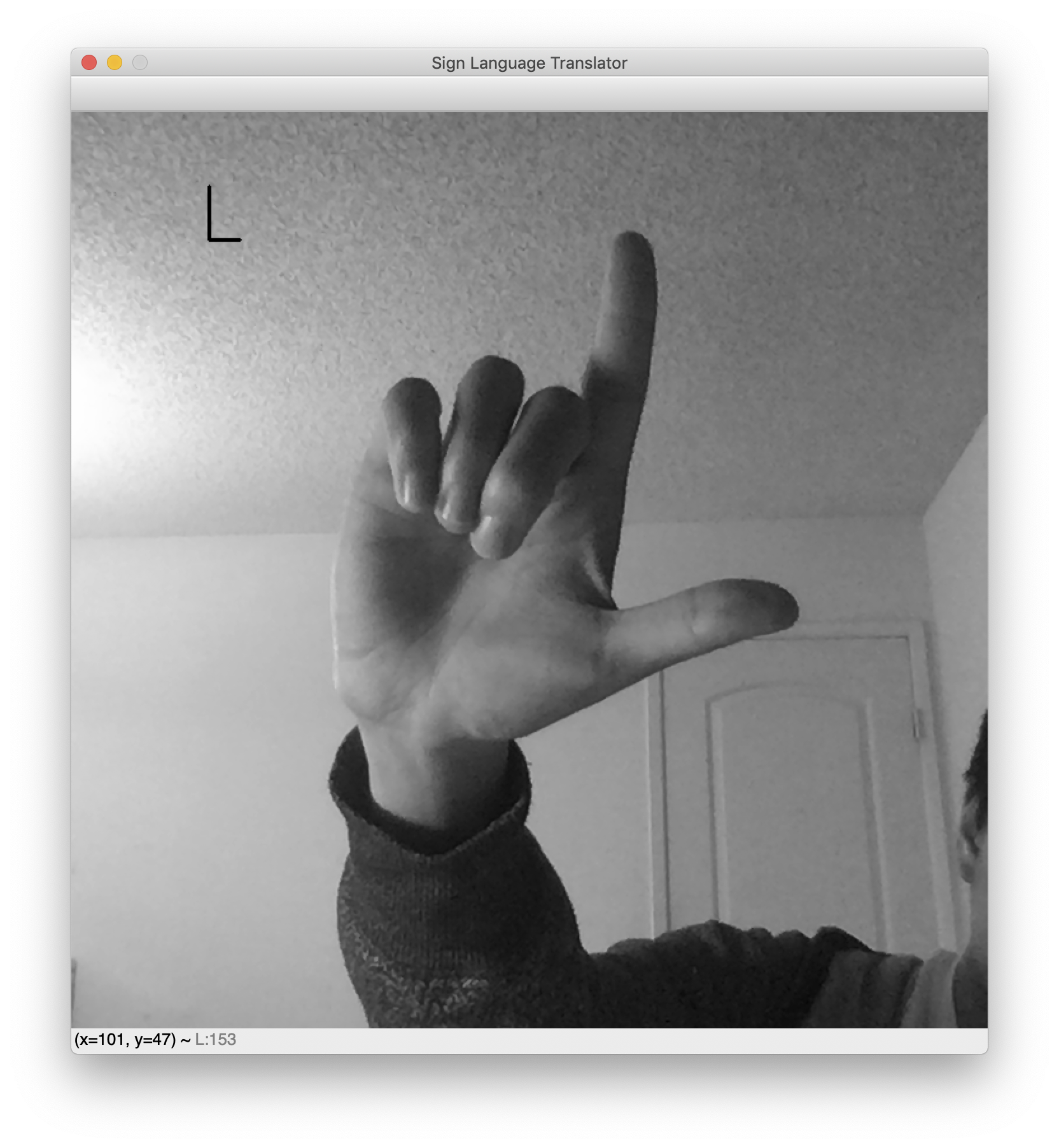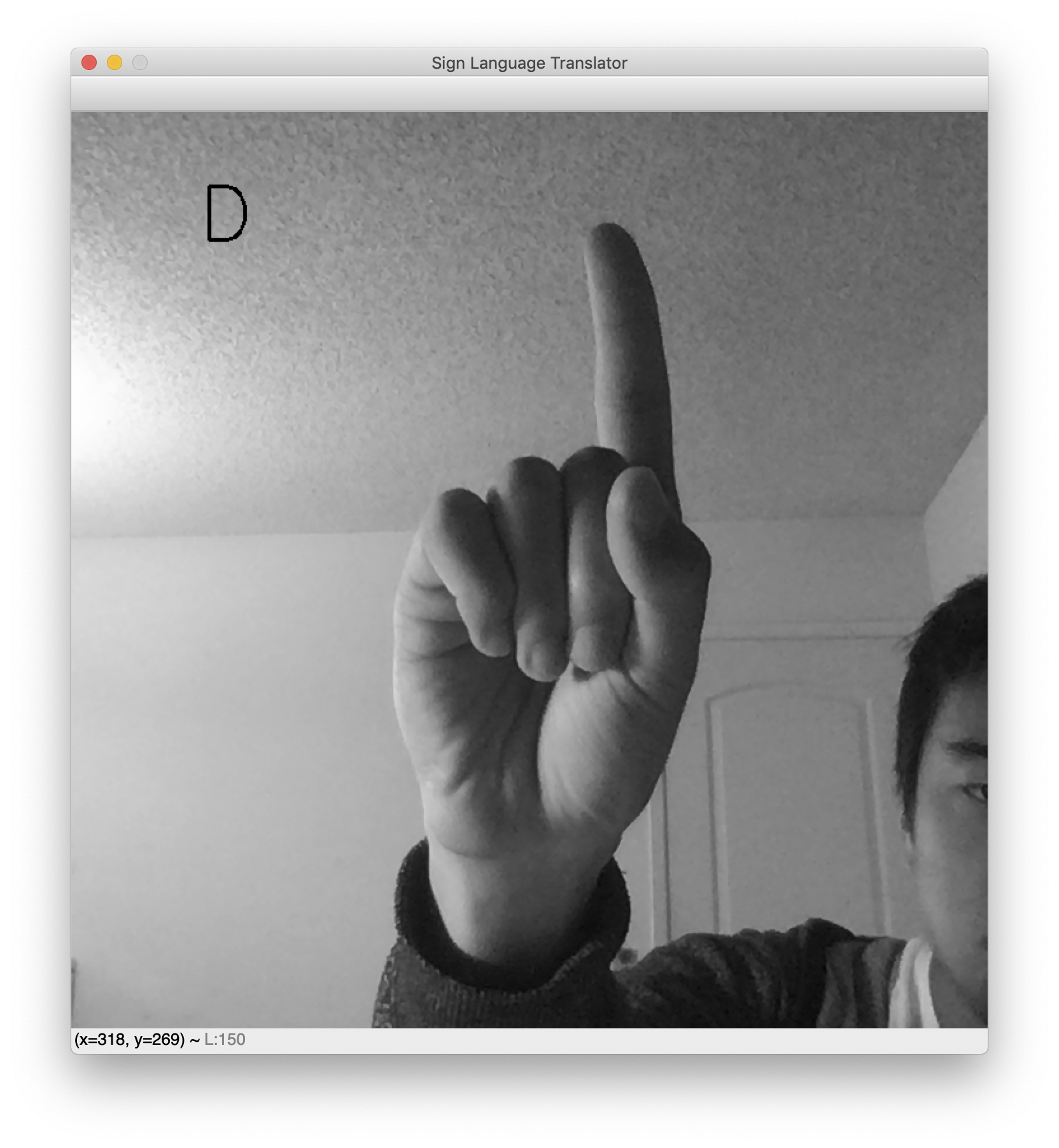While testing, note that the background needs to be fairly clear for this translator to work. This is an unfortunate consequence of the dataset’s cleanliness. Had the dataset included images of hand signs with miscellaneous backgrounds, the network would be robust to noisy backgrounds. However, the dataset features blank backgrounds and nicely centered hands. As a result, this webcam translator works best when your hand is likewise centered and placed against a blank background.

This concludes the sign language translator application.

## Conclusion

In this tutorial, you built an American Sign Language translator using computer vision and a machine learning model. In particular, you saw new aspects of training a machine learning model—specifically, data augmentation for model robustness, learning rate schedules for lower loss, and exporting AI models using ONNX for production use. This then culminated in a real-time computer vision application, which translates sign language into letters using a pipeline you built. It’s worth noting that combatting the brittleness of the final classifier can be tackled with any or all of the following methods. For further exploration try the following topics to in improve your application:

• Generalization: This isn’t a sub-topic within computer vision, rather, it’s a constant problem throughout all of machine learning. See Understanding Bias-Variance Tradeoffs.
• Domain Adaptation: Say your model is trained in domain A (for example, sunny environments). Can you adapt the model to domain B (for example, cloudy environments) quickly?
• Adversarial Examples: Say an adversary is designing images intentionally to fool your model. How can you design such images? How can you combat such images?
]]>
Alvin Wan 2020-05-12T20:33:31.626Z
<![CDATA[Installing "pcalg" on RStudio]]> tag:www.digitalocean.com,2005:/community/questions/installing-pcalg-on-rstudio 2019-07-12T14:02:11.263Z Dear community,

I’m new to DigitalOcean and still lack experience. I set up a Droplet with RStudio on Ubuntu 18.04. To run my code, I need to install the “pcalg” package. However, I always get the following error messsage. Does anyone have an idea how to fix this? Your help would be much appreciated.

``````> install.packages("pcalg")
Installing package into ‘/home/paul/R/x86_64-pc-linux-gnu-library/3.6’
(as ‘lib’ is unspecified)
Warning in install.packages :
dependencies ‘graph’, ‘RBGL’ are not available
also installing the dependencies ‘V8’, ‘dagitty’

trying URL 'https://cloud.r-project.org/src/contrib/V8_2.3.tar.gz'
Content type 'application/x-gzip' length 304765 bytes (297 KB)
==================================================

trying URL 'https://cloud.r-project.org/src/contrib/dagitty_0.2-2.tar.gz'
Content type 'application/x-gzip' length 168082 bytes (164 KB)
==================================================

trying URL 'https://cloud.r-project.org/src/contrib/pcalg_2.6-2.tar.gz'
Content type 'application/x-gzip' length 4859003 bytes (4.6 MB)
==================================================

* installing *source* package ‘V8’ ...
** package ‘V8’ successfully unpacked and MD5 sums checked
** using staged installation
Using PKG_CFLAGS=-I/usr/include/v8 -I/usr/include/v8-3.14
Using PKG_LIBS=-lv8 -lv8_libplatform
------------------------- ANTICONF ERROR ---------------------------
* deb: libv8-dev or libnode-dev (Debian / Ubuntu)
* rpm: v8-devel (Fedora, EPEL)
* brew: v8 (OSX)
* csw: libv8_dev (Solaris)
To use a custom libv8, set INCLUDE_DIR and LIB_DIR manually via:
R CMD INSTALL --configure-vars='INCLUDE_DIR=... LIB_DIR=...'
--------------------------------------------------------------------
ERROR: configuration failed for package ‘V8’
* removing ‘/home/paul/R/x86_64-pc-linux-gnu-library/3.6/V8’
Warning in install.packages :
installation of package ‘V8’ had non-zero exit status
ERROR: dependency ‘V8’ is not available for package ‘dagitty’
* removing ‘/home/paul/R/x86_64-pc-linux-gnu-library/3.6/dagitty’
Warning in install.packages :
installation of package ‘dagitty’ had non-zero exit status
ERROR: dependencies ‘graph’, ‘RBGL’, ‘dagitty’ are not available for package ‘pcalg’
* removing ‘/home/paul/R/x86_64-pc-linux-gnu-library/3.6/pcalg’
Warning in install.packages :
installation of package ‘pcalg’ had non-zero exit status

``````
]]>
PHU 2019-07-12T14:02:11.263Z
<![CDATA[Python Machine Learning Projects — A DigitalOcean eBook]]> tag:www.digitalocean.com,2005:/community/tutorials/python-machine-learning-projects-a-digitalocean-ebook 2019-05-02T16:59:07.248Z

Machine Learning Projects: Python eBook in EPUB format

Machine Learning Projects: Python eBook in PDF format

Machine Learning Projects: Python eBook in Mobi format

## Introduction to the eBook

As machine learning is increasingly leveraged to find patterns, conduct analysis, and make decisions — sometimes without final input from humans who may be impacted by these findings — it is crucial to invest in bringing more stakeholders into the fold. This book of Python projects in machine learning tries to do just that: to equip the developers of today and tomorrow with tools they can use to better understand, evaluate, and shape machine learning to help ensure that it is serving us all.

This book will set you up with a Python programming environment if you don’t have one already, then provide you with a conceptual understanding of machine learning in the chapter “An Introduction to Machine Learning.” What follows next are three Python machine learning projects. They will help you create a machine learning classifier, build a neural network to recognize handwritten digits, and give you a background in deep reinforcement learning through building a bot for Atari.

These chapters originally appeared as articles on DigitalOcean Community, written by members of the international software developer community. If you are interested in contributing to this knowledge base, consider proposing a tutorial to the Write for DOnations program. DigitalOcean offers payment to authors and provides a matching donation to tech-focused nonprofits.

## Other Books in this Series

If you are learning Python or are looking for reference material, you can download our free Python eBook, How To Code in Python 3

For other programming languages and DevOps engineering articles, check out our knowledge base of over 2,100 tutorials.

You can download the eBook in either the EPUB, PDF, or Mobi format by following the links below.

]]>
Lisa Tagliaferri Brian Boucheron 2019-05-02T16:59:07.248Z
<![CDATA[How To Apply Computer Vision to Build an Emotion-Based Dog Filter in Python 3]]> tag:www.digitalocean.com,2005:/community/tutorials/how-to-apply-computer-vision-to-build-an-emotion-based-dog-filter-in-python-3 2019-04-03T19:18:50.082Z The author selected Girls Who Code to receive a donation as part of the Write for DOnations program.

### Introduction

Computer vision is a subfield of computer science that aims to extract a higher-order understanding from images and videos. This field includes tasks such as object detection, image restoration (matrix completion), and optical flow. Computer vision powers technologies such as self-driving car prototypes, employee-less grocery stores, fun Snapchat filters, and your mobile device’s face authenticator.

In this tutorial, you will explore computer vision as you use pre-trained models to build a Snapchat-esque dog filter. For those unfamiliar with Snapchat, this filter will detect your face and then superimpose a dog mask on it. You will then train a face-emotion classifier so that the filter can pick dog masks based on emotion, such as a corgi for happy or a pug for sad. Along the way, you will also explore related concepts in both ordinary least squares and computer vision, which will expose you to the fundamentals of machine learning.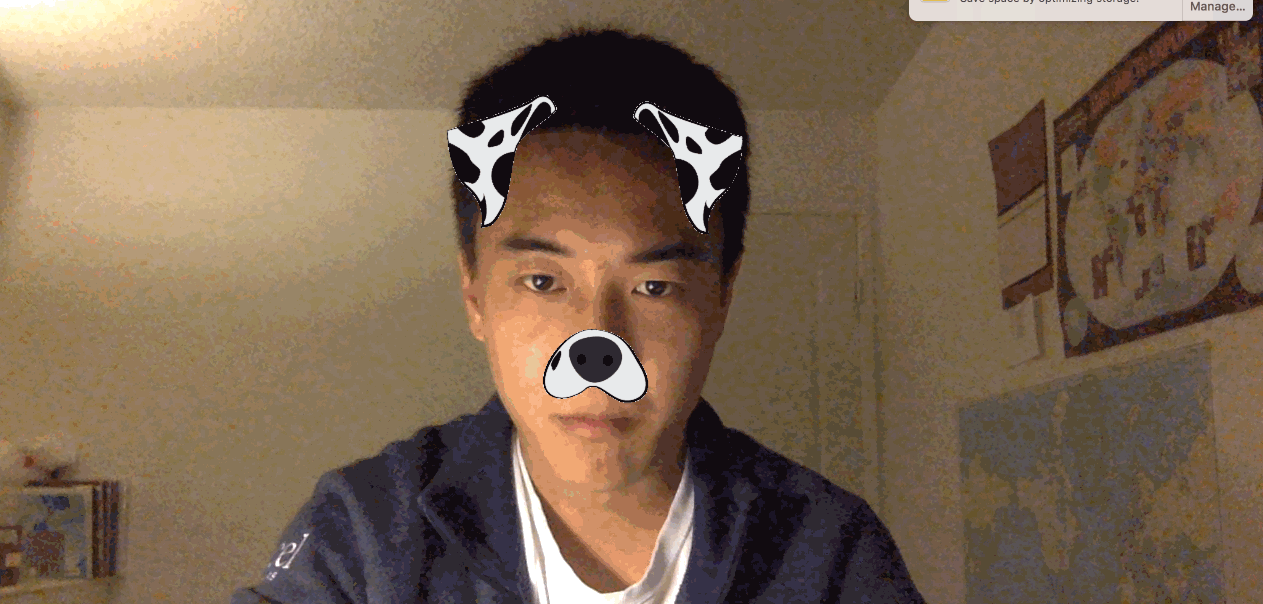As you work through the tutorial, you’ll use `OpenCV`, a computer-vision library, `numpy` for linear algebra utilities, and `matplotlib` for plotting. You’ll also apply the following concepts as you build a computer-vision application:

• Ordinary least squares as a regression and classification technique.
• The basics of stochastic gradient neural networks.

While not necessary to complete this tutorial, you’ll find it easier to understand some of the more detailed explanations if you’re familiar with these mathematical concepts:

• Fundamental linear algebra concepts: scalars, vectors, and matrices.
• Fundamental calculus: how to take a derivative.

You can find the complete code for this tutorial at https://github.com/do-community/emotion-based-dog-filter.

Let’s get started.

## Prerequisites

To complete this tutorial, you will need the following:

## Step 1 — Creating The Project and Installing Dependencies

Let’s create a workspace for this project and install the dependencies we’ll need. We’ll call our workspace `DogFilter`:

``````mkdir ~/DogFilter

``````

Navigate to the `DogFilter` directory:

``````cd ~/DogFilter

``````

Then create a new Python virtual environment for the project:

``````python3 -m venv dogfilter

``````

``````source dogfilter/bin/activate

``````

The prompt changes, indicating the environment is active. Now install PyTorch, a deep-learning framework for Python that we’ll use in this tutorial. The installation process depends on which operating system you’re using.

On macOS, install Pytorch with the following command:

``````python -m pip install torch==0.4.1 torchvision==0.2.1

``````

On Linux, use the following commands:

``````pip install http://download.pytorch.org/whl/cpu/torch-0.4.1-cp35-cp35m-linux_x86_64.whl
pip install torchvision

``````

And for Windows, install Pytorch with these commands:

``````pip install http://download.pytorch.org/whl/cpu/torch-0.4.1-cp35-cp35m-win_amd64.whl
pip install torchvision

``````

Now install prepackaged binaries for `OpenCV` and `numpy`, which are computer vision and linear algebra libraries, respectively. The former offers utilities such as image rotations, and the latter offers linear algebra utilities such as a matrix inversion.

``````python -m pip install opencv-python==3.4.3.18 numpy==1.14.5

``````

Finally, create a directory for our assets, which will hold the images we’ll use in this tutorial:

``````mkdir assets

``````

With the dependencies installed, let’s build the first version of our filter: a face detector.

## Step 2 — Building a Face Detector

Our first objective is to detect all faces in an image. We’ll create a script that accepts a single image and outputs an annotated image with the faces outlined with boxes.

Fortunately, instead of writing our own face detection logic, we can use pre-trained models. We’ll set up a model and then load pre-trained parameters. OpenCV makes this easy by providing both.

OpenCV provides the model parameters in its source code. but we need the absolute path to our locally-installed OpenCV to use these parameters. Since that absolute path may vary, we’ll download our own copy instead and place it in the `assets` folder:

``````wget -O assets/haarcascade_frontalface_default.xml https://github.com/opencv/opencv/raw/master/data/haarcascades/haarcascade_frontalface_default.xml

``````

The `-O` option specifies the destination as `assets/haarcascade_frontalface_default.xml`. The second argument is the source URL.

We’ll detect all faces in the following image from Pexels (CC0, link to original image).First, download the image. The following command saves the downloaded image as `children.png` in the `assets` folder:

``````wget -O assets/children.png https://assets.digitalocean.com/articles/python3_dogfilter/CfoBWbF.png

``````

To check that the detection algorithm works, we will run it on an individual image and save the resulting annotated image to disk. Create an `outputs` folder for these annotated results.

``````mkdir outputs

``````

Now create a Python script for the face detector. Create the file `step_1_face_detect` using `nano` or your favorite text editor:

``````nano step_2_face_detect.py

``````

Add the following code to the file. This code imports OpenCV, which contains the image utilities and face classifier. The rest of the code is typical Python program boilerplate.

step_2_face_detect.py
``````"""Test for face detection"""

import cv2

def main():
pass

if __name__ == '__main__':
main()
``````

Now replace `pass` in the `main` function with this code which initializes a face classifier using the OpenCV parameters you downloaded to your `assets` folder:

step_2_face_detect.py
``````def main():
# initialize front face classifier
``````

Next, add this line to load the image `children.png`.

step_2_face_detect.py
``````    frame = cv2.imread('assets/children.png')
``````

Then add this code to convert the image to black and white, as the classifier was trained on black-and-white images. To accomplish this, we convert to grayscale and then discretize the histogram:

step_2_face_detect.py
``````    # Convert to black-and-white
gray = cv2.cvtColor(frame, cv2.COLOR_BGR2GRAY)
blackwhite = cv2.equalizeHist(gray)
``````

Then use OpenCV’s `detectMultiScale` function to detect all faces in the image.

step_2_face_detect.py
``````    rects = cascade.detectMultiScale(
blackwhite, scaleFactor=1.3, minNeighbors=4, minSize=(30, 30),
``````
• `scaleFactor` specifies how much the image is reduced along each dimension.
• `minNeighbors` denotes how many neighboring rectangles a candidate rectangle needs to be retained.
• `minSize` is the minimum allowable detected object size. Objects smaller than this are discarded.

The return type is a list of tuples, where each tuple has four numbers denoting the minimum x, minimum y, width, and height of the rectangle in that order.

Iterate over all detected objects and draw them on the image in green using `cv2.rectangle`:

step_2_face_detect.py
``````    for x, y, w, h in rects:
cv2.rectangle(frame, (x, y), (x + w, y + h), (0, 255, 0), 2)
``````
• The second and third arguments are opposing corners of the rectangle.
• The fourth argument is the color to use. `(0, 255, 0)` corresponds to green for our RGB color space.
• The last argument denotes the width of our line.

Finally, write the image with bounding boxes into a new file at `outputs/children_detected.png`:

step_2_face_detect.py
``````    cv2.imwrite('outputs/children_detected.png', frame)
``````

Your completed script should look like this:

step_2_face_detect.py
``````"""Tests face detection for a static image."""

import cv2

def main():

# initialize front face classifier

# Convert to black-and-white
gray = cv2.cvtColor(frame, cv2.COLOR_BGR2GRAY)
blackwhite = cv2.equalizeHist(gray)

blackwhite, scaleFactor=1.3, minNeighbors=4, minSize=(30, 30),

for x, y, w, h in rects:
cv2.rectangle(frame, (x, y), (x + w, y + h), (0, 255, 0), 2)

cv2.imwrite('outputs/children_detected.png', frame)

if __name__ == '__main__':
main()
``````

Save the file and exit your editor. Then run the script:

``````python step_2_face_detect.py

``````

Open `outputs/children_detected.png`. You’ll see the following image that shows the faces outlined with boxes: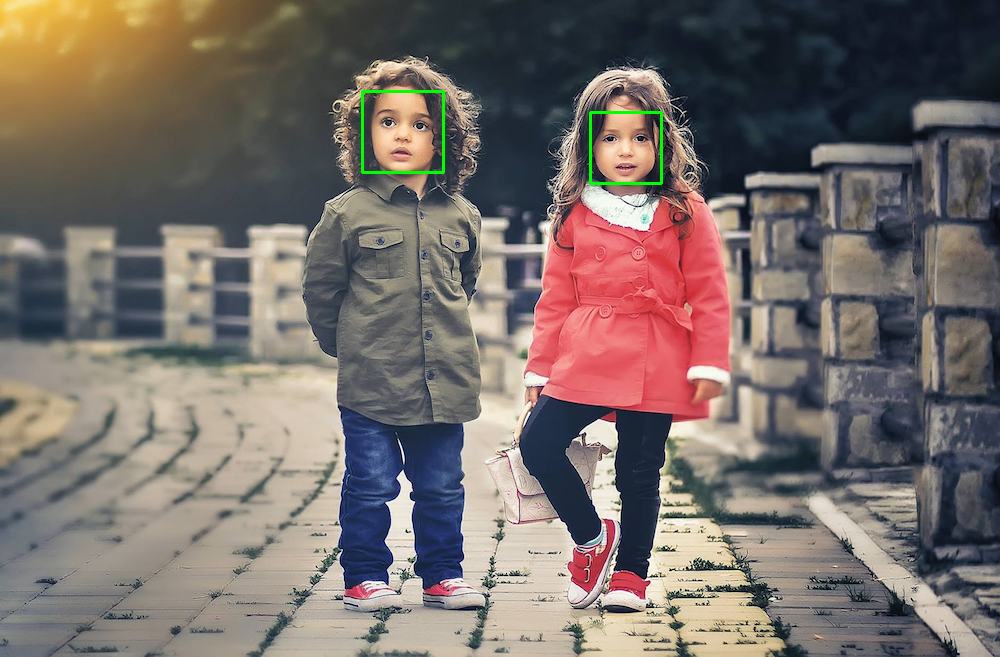At this point, you have a working face detector. It accepts an image as input and draws bounding boxes around all faces in the image, outputting the annotated image. Now let’s apply this same detection to a live camera feed.

## Step 3 — Linking the Camera Feed

The next objective is to link the computer’s camera to the face detector. Instead of detecting faces in a static image, you’ll detect all faces from your computer’s camera. You will collect camera input, detect and annotate all faces, and then display the annotated image back to the user. You’ll continue from the script in Step 2, so start by duplicating that script:

``````cp step_2_face_detect.py step_3_camera_face_detect.py

``````

Then open the new script in your editor:

``````nano step_3_camera_face_detect.py

``````

You will update the `main` function by using some elements from this test script from the official OpenCV documentation. Start by initializing a `VideoCapture` object that is set to capture live feed from your computer’s camera. Place this at the start of the `main` function, before the other code in the function:

step_3_camera_face_detect.py
``````def main():
cap = cv2.VideoCapture(0)
...
``````

Starting from the line defining `frame`, indent all of your existing code, placing all of the code in a `while` loop.

step_3_camera_face_detect.py
``````    while True:
...
for x, y, w, h in rects:
cv2.rectangle(frame, (x, y), (x + w, y + h), (0, 255, 0), 2)

cv2.imwrite('outputs/children_detected.png', frame)
``````

Replace the line defining `frame` at the start of the `while` loop. Instead of reading from an image on disk, you’re now reading from the camera:

step_3_camera_face_detect.py
``````    while True:
# frame = cv2.imread('assets/children.png') # DELETE ME
# Capture frame-by-frame
``````

Replace the line `cv2.imwrite(...)` at the end of the `while` loop. Instead of writing an image to disk, you’ll display the annotated image back to the user’s screen:

step_3_camera_face_detect.py
``````      cv2.imwrite('outputs/children_detected.png', frame)  # DELETE ME
# Display the resulting frame
cv2.imshow('frame', frame)
``````

Also, add some code to watch for keyboard input so you can stop the program. Check if the user hits the `q` character and, if so, quit the application. Right after `cv2.imshow(...)` add the following:

step_3_camera_face_detect.py
``````...
cv2.imshow('frame', frame)
if cv2.waitKey(1) & 0xFF == ord('q'):
break
...
``````

The line `cv2.waitkey(1)` halts the program for 1 millisecond so that the captured image can be displayed back to the user.

Finally, release the capture and close all windows. Place this outside of the `while` loop to end the `main` function.

step_3_camera_face_detect.py
``````...

while True:
...

cap.release()
cv2.destroyAllWindows()
``````

Your script should look like the following:

step_3_camera_face_detect.py
``````"""Test for face detection on video camera.

Move your face around and a green box will identify your face.
With the test frame in focus, hit `q` to exit.
Note that typing `q` into your terminal will do nothing.
"""

import cv2

def main():
cap = cv2.VideoCapture(0)

# initialize front face classifier

while True:
# Capture frame-by-frame

# Convert to black-and-white
gray = cv2.cvtColor(frame, cv2.COLOR_BGR2GRAY)
blackwhite = cv2.equalizeHist(gray)

# Detect faces
blackwhite, scaleFactor=1.3, minNeighbors=4, minSize=(30, 30),

# Add all bounding boxes to the image
for x, y, w, h in rects:
cv2.rectangle(frame, (x, y), (x + w, y + h), (0, 255, 0), 2)

# Display the resulting frame
cv2.imshow('frame', frame)
if cv2.waitKey(1) & 0xFF == ord('q'):
break

# When everything done, release the capture
cap.release()
cv2.destroyAllWindows()

if __name__ == '__main__':
main()
``````

Save the file and exit your editor.

Now run the test script.

``````python step_3_camera_face_detect.py

``````

This activates your camera and opens a window displaying your camera’s feed. Your face will be boxed by a green square in real time: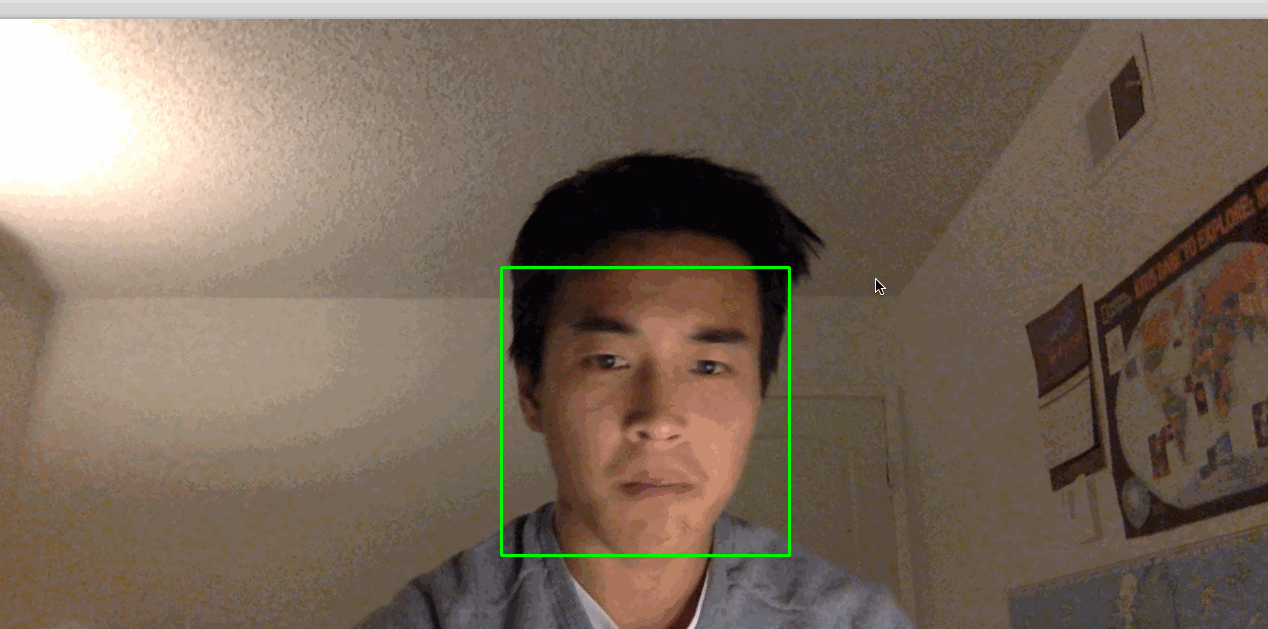Note: If you find that you have to hold very still for things to work, the lighting in the room may not be adequate. Try moving to a brightly lit room where you and your background have high constrast. Also, avoid bright lights near your head. For example, if you have your back to the sun, this process might not work very well.

Our next objective is to take the detected faces and superimpose dog masks on each one.

## Step 4 — Building the Dog Filter

Before we build the filter itself, let’s explore how images are represented numerically. This will give you the background needed to modify images and ultimately apply a dog filter.

Let’s look at an example. We can construct a black-and-white image using numbers, where `0` corresponds to black and `1` corresponds to white.

Focus on the dividing line between 1s and 0s. What shape do you see?

``````0 0 0 0 0 0 0 0 0
0 0 0 0 1 0 0 0 0
0 0 0 1 1 1 0 0 0
0 0 1 1 1 1 1 0 0
0 0 0 1 1 1 0 0 0
0 0 0 0 1 0 0 0 0
0 0 0 0 0 0 0 0 0
``````

The image is a diamond. If save this matrix of values as an image. This gives us the following picture:We can use any value between 0 and 1, such as 0.1, 0.26, or 0.74391. Numbers closer to 0 are darker and numbers closer to 1 are lighter. This allows us to represent white, black, and any shade of gray. This is great news for us because we can now construct any grayscale image using 0, 1, and any value in between. Consider the following, for example. Can you tell what it is? Again, each number corresponds to the color of a pixel.

``````1  1  1  1  1  1  1  1  1  1  1  1
1  1  1  1  0  0  0  0  1  1  1  1
1  1  0  0 .4 .4 .4 .4  0  0  1  1
1  0 .4 .4 .5 .4 .4 .4 .4 .4  0  1
1  0 .4 .5 .5 .5 .4 .4 .4 .4  0  1
0 .4 .4 .4 .5 .4 .4 .4 .4 .4 .4  0
0 .4 .4 .4 .4  0  0 .4 .4 .4 .4  0
0  0 .4 .4  0  1 .7  0 .4 .4  0  0
0  1  0  0  0 .7 .7  0  0  0  1  0
1  0  1  1  1  0  0 .7 .7 .4  0  1
1  0 .7  1  1  1 .7 .7 .7 .7  0  1
1  1  0  0 .7 .7 .7 .7  0  0  1  1
1  1  1  1  0  0  0  0  1  1  1  1
1  1  1  1  1  1  1  1  1  1  1  1
``````

Re-rendered as an image, you can now tell that this is, in fact, a Poké Ball:You’ve now seen how black-and-white and grayscale images are represented numerically. To introduce color, we need a way to encode more information. An image has its height and width expressed as `h x w`.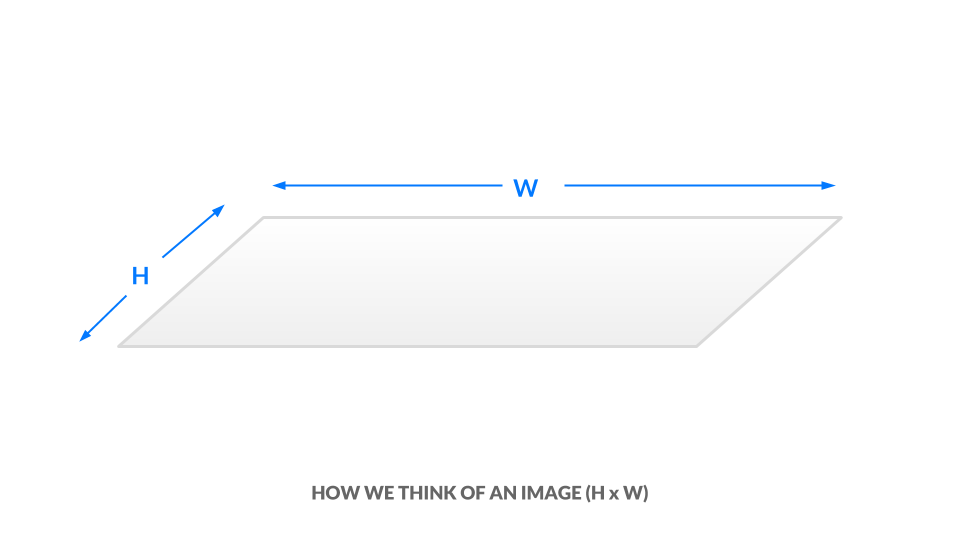In the current grayscale representation, each pixel is one value between 0 and 1. We can equivalently say our image has dimensions `h x w x 1`. In other words, every `(x, y)` position in our image has just one value.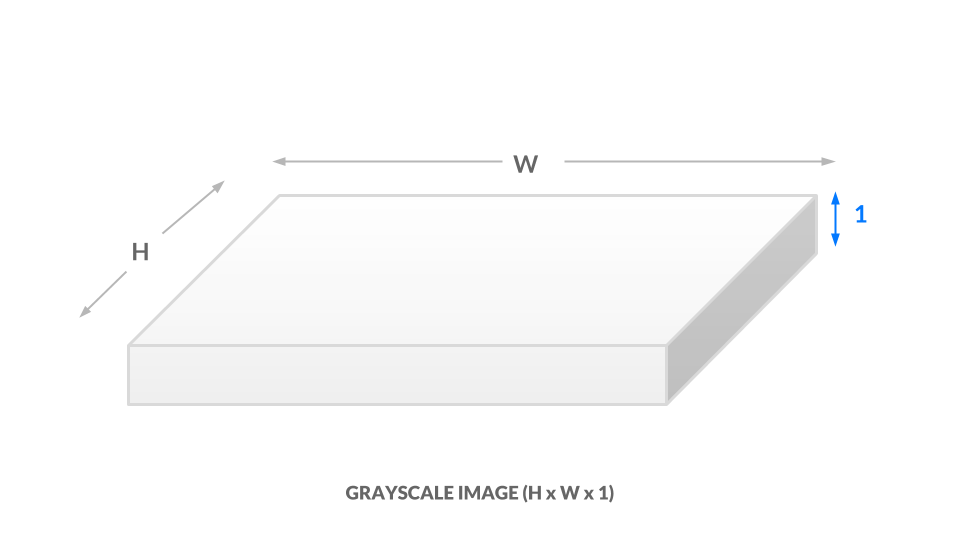For a color representation, we represent the color of each pixel using three values between 0 and 1. One number corresponds to the “degree of red,” one to the “degree of green,” and the last to the “degree of blue.” We call this the RGB color space. This means that for every `(x, y)` position in our image, we have three values `(r, g, b)`. As a result, our image is now `h x w x 3`: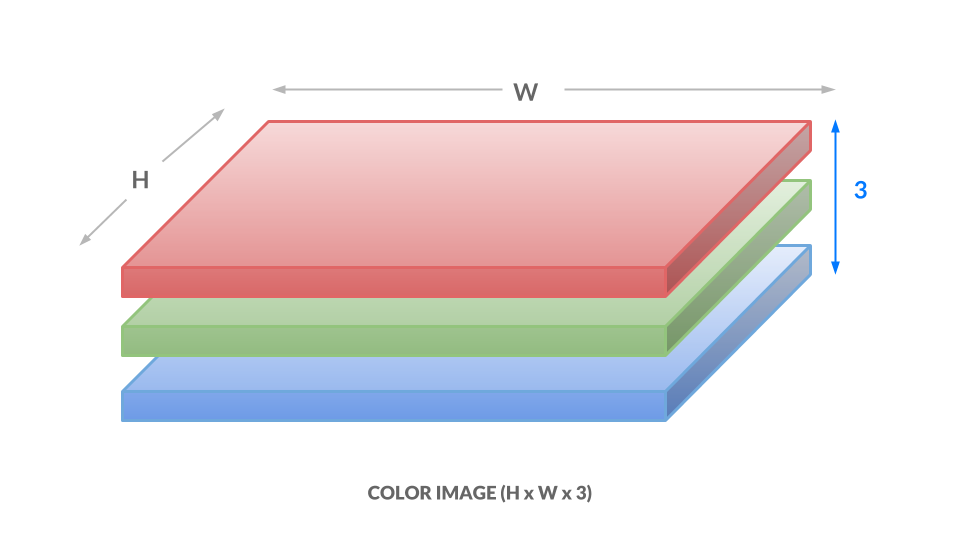Here, each number ranges from 0 to 255 instead of 0 to 1, but the idea is the same. Different combinations of numbers correspond to different colors, such as dark purple `(102, 0, 204)` or bright orange `(255, 153, 51)`. The takeaways are as follows:

1. Each image will be represented as a box of numbers that has three dimensions: height, width, and color channels. Manipulating this box of numbers directly is equivalent to manipulating the image.
2. We can also flatten this box to become just a list of numbers. In this way, our image becomes a vector. Later on, we will refer to images as vectors.

Now that you understand how images are represented numerically, you are well-equipped to begin applying dog masks to faces. To apply a dog mask, you will replace values in the child image with non-white dog mask pixels. To start, you will work with a single image. Download this crop of a face from the image you used in Step 2.

``````wget -O assets/child.png https://assets.digitalocean.com/articles/python3_dogfilter/alXjNK1.png

``````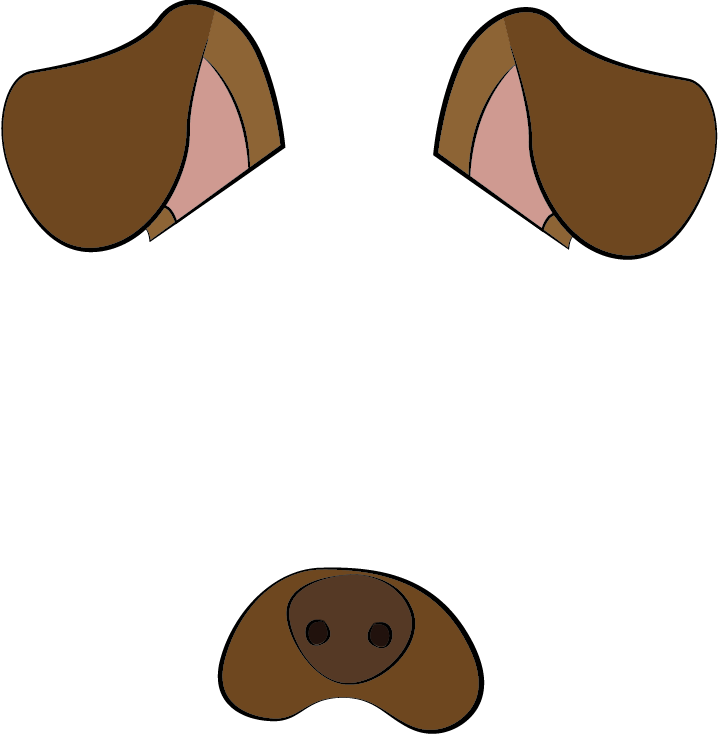Download this with `wget`:

``````wget -O assets/dog.png https://assets.digitalocean.com/articles/python3_dogfilter/ED32BCs.png

``````

Create a new file called `step_4_dog_mask_simple.py` which will hold the code for the script that applies the dog mask to faces:

``````nano step_4_dog_mask_simple.py

``````

Add the following boilerplate for the Python script and import the OpenCV and `numpy` libraries:

``````"""Test for adding dog mask"""

import cv2
import numpy as np

def main():
pass

if __name__ == '__main__':
main()
``````

Replace `pass` in the `main` function with these two lines which load the original image and the dog mask into memory.

``````...
def main():
``````

Next, fit the dog mask to the child. The logic is more complicated than what we’ve done previously, so we will create a new function called `apply_mask` to modularize our code. Directly after the two lines that load the images, add this line which invokes the `apply_mask` function:

``````...
``````

Create a new function called `apply_mask` and place it above the `main` function:

``````...
pass

def main():
...
``````

At this point, your file should look like this:

``````"""Test for adding dog mask"""

import cv2
import numpy as np

pass

def main():

if __name__ == '__main__':
main()
``````

Let’s build out the `apply_mask` function. Our goal is to apply the mask to the child’s face. However, we need to maintain the aspect ratio for our dog mask. To do so, we need to explicitly compute our dog mask’s final dimensions. Inside the `apply_mask` function, replace `pass` with these two lines which extract the height and width of both images:

``````...
face_h, face_w, _ = face.shape
``````

Next, determine which dimension needs to be “shrunk more.” To be precise, we need the tighter of the two constraints. Add this line to the `apply_mask` function:

``````...

# Resize the mask to fit on face
``````

Then compute the new shape by adding this code to the function:

``````...
``````

Here we cast the numbers to integers, as the `resize` function needs integral dimensions.

Now add this code to resize the dog mask to the new shape:

``````...

``````

Finally, write the image to disk so you can double-check that your resized dog mask is correct after you run the script:

``````    cv2.imwrite('outputs/resized_dog.png', resized_mask)
``````

The completed script should look like this:

``````"""Test for adding dog mask"""
import cv2
import numpy as np

face_h, face_w, _ = face.shape

# Resize the mask to fit on face

def main():

if __name__ == '__main__':
main()

``````

Save the file and exit your editor. Run the new script:

``````python step_4_dog_mask_simple.py

``````

Open the image at `outputs/resized_dog.png` to double-check the mask was resized correctly. It will match the dog mask shown earlier in this section.

Now add the dog mask to the child. Open the `step_4_dog_mask_simple.py` file again and return to the `apply_mask` function:

``````nano step_4_dog_mask_simple.py

``````

First, remove the line of code that writes the resized mask from the `apply_mask` function since you no longer need it:

``````    cv2.imwrite('outputs/resized_dog.png', resized_mask)  # delete this line
...
``````

In its place, apply your knowledge of image representation from the start of this section to modify the image. Start by making a copy of the child image. Add this line to the `apply_mask` function:

``````...
``````

Next, find all positions where the dog mask is not white or near white. To do this, check if the pixel value is less than 250 across all color channels, as we’d expect a near-white pixel to be near `[255, 255, 255]`. Add this code:

``````...
``````

At this point, the dog image is, at most, as large as the child image. We want to center the dog image on the face, so compute the offset needed to center the dog image by adding this code to `apply_mask`:

``````...
off_h = int((face_h - new_mask_h) / 2)
off_w = int((face_w - new_mask_w) / 2)
``````

Copy all non-white pixels from the dog image into the child image. Since the child image may be larger than the dog image, we need to take a subset of the child image:

``````    face_with_mask[off_h: off_h+new_mask_h, off_w: off_w+new_mask_w][non_white_pixels] = \
``````

Then return the result:

``````    return face_with_mask
``````

In the `main` function, add this code to write the result of the `apply_mask` function to an output image so you can manually double-check the result:

``````...
``````

Your completed script will look like the following:

``````"""Test for adding dog mask"""

import cv2
import numpy as np

face_h, face_w, _ = face.shape

# Resize the mask to fit on face

off_h = int((face_h - new_mask_h) / 2)
off_w = int((face_w - new_mask_w) / 2)

def main():

if __name__ == '__main__':
main()
``````

Save the script and run it:

``````python step_4_dog_mask_simple.py

``````

You’ll have the following picture of a child with a dog mask in `outputs/child_with_dog_mask.png`:You now have a utility that applies dog masks to faces. Now let’s use what you’ve built to add the dog mask in real time.

We’ll pick up from where we left off in Step 3. Copy `step_3_camera_face_detect.py` to `step_4_dog_mask.py`.

``````cp step_3_camera_face_detect.py step_4_dog_mask.py

``````

``````nano step_4_dog_mask.py

``````

First, import the NumPy library at the top of the script:

``````import numpy as np
...
``````

Then add the `apply_mask` function from your previous work into this new file above the `main` function:

``````def apply_mask(face: np.array, mask: np.array) -> np.array:
face_h, face_w, _ = face.shape

# Resize the mask to fit on face

off_h = int((face_h - new_mask_h) / 2)
off_w = int((face_w - new_mask_w) / 2)

...
``````

Second, locate this line in the `main` function:

``````    cap = cv2.VideoCapture(0)
``````

``````    cap = cv2.VideoCapture(0)

...
``````

Next, in the `while` loop, locate this line:

``````        ret, frame = cap.read()
``````

Add this line after it to extract the image’s height and width:

``````        ret, frame = cap.read()
frame_h, frame_w, _ = frame.shape
...
``````

Next, delete the line in `main` that draws bounding boxes. You’ll find this line in the `for` loop that iterates over detected faces:

``````        for x, y, w, h in rects:
...
cv2.rectangle(frame, (x, y), (x + w, y + h), (0, 255, 0), 2) # DELETE ME
...
``````

In its place, add this code which crops the frame. For aesthetic purposes, we crop an area slightly larger than the face.

``````        for x, y, w, h in rects:
# crop a frame slightly larger than the face
y0, y1 = int(y - 0.25*h), int(y + 0.75*h)
x0, x1 = x, x + w
``````

Introduce a check in case the detected face is too close to the edge.

``````            # give up if the cropped frame would be out-of-bounds
if x0 < 0 or y0 < 0 or x1 > frame_w or y1 > frame_h:
continue
``````

Finally, insert the face with a mask into the image.

``````            # apply mask
``````

Verify that your script looks like this:

``````"""Real-time dog filter

Move your face around and a dog filter will be applied to your face if it is not out-of-bounds. With the test frame in focus, hit `q` to exit. Note that typing `q` into your terminal will do nothing.
"""

import numpy as np
import cv2

face_h, face_w, _ = face.shape

# Resize the mask to fit on face

off_h = int((face_h - new_mask_h) / 2)
off_w = int((face_w - new_mask_w) / 2)

def main():
cap = cv2.VideoCapture(0)

# initialize front face classifier

while(True):
# Capture frame-by-frame
frame_h, frame_w, _ = frame.shape

# Convert to black-and-white
gray = cv2.cvtColor(frame, cv2.COLOR_BGR2GRAY)
blackwhite = cv2.equalizeHist(gray)

# Detect faces
blackwhite, scaleFactor=1.3, minNeighbors=4, minSize=(30, 30),

for x, y, w, h in rects:
# crop a frame slightly larger than the face
y0, y1 = int(y - 0.25*h), int(y + 0.75*h)
x0, x1 = x, x + w

# give up if the cropped frame would be out-of-bounds
if x0 < 0 or y0 < 0 or x1 > frame_w or y1 > frame_h:
continue

# Display the resulting frame
cv2.imshow('frame', frame)
if cv2.waitKey(1) & 0xFF == ord('q'):
break

# When everything done, release the capture
cap.release()
cv2.destroyAllWindows()

if __name__ == '__main__':
main()
``````

Save the file and exit your editor. Then run the script.

``````python step_4_dog_mask.py

``````

You now have a real-time dog filter running. The script will also work with multiple faces in the picture, so you can get your friends together for some automatic dog-ification.This concludes our first primary objective in this tutorial, which is to create a Snapchat-esque dog filter. Now let’s use facial expression to determine the dog mask applied to a face.

## Step 5 — Build a Basic Face Emotion Classifier using Least Squares

In this section you’ll create an emotion classifier to apply different masks based on displayed emotions. If you smile, the filter will apply a corgi mask. If you frown, it will apply a pug mask. Along the way, you’ll explore the least-squares framework, which is fundamental to understanding and discussing machine learning concepts.

To understand how to process our data and produce predictions, we’ll first briefly explore machine learning models.

We need to ask two questions for each model that we consider. For now, these two questions will be sufficient to differentiate between models:

1. Input: What information is the model given?
2. Output: What is the model trying to predict?

At a high-level, the goal is to develop a model for emotion classification. The model is:

1. Input: given images of faces.
2. Output: predicts the corresponding emotion.
``````model: face -> emotion
``````

The approach we’ll use is least squares; we take a set of points, and we find a line of best fit. The line of best fit, shown in the following image, is our model.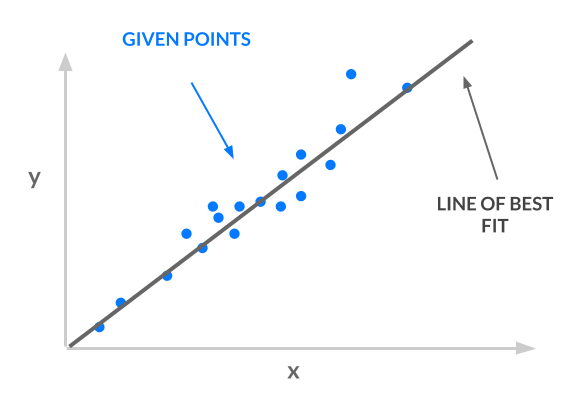Consider the input and output for our line:

1. Input: given `x` coordinates.
2. Output: predicts the corresponding \$y\$ coordinate.
``````least squares line: x -> y
``````

Our input `x` must represent faces and our output `y` must represent emotion, in order for us to use least squares for emotion classification:

• `x -> face`: Instead of using one number for `x`, we will use a vector of values for `x`. Thus, `x` can represent images of faces. The article Ordinary Least Squares explains why you can use a vector of values for `x`.
• `y -> emotion`: Each emotion will correspond to a number. For example, “angry” is 0, “sad” is 1, and “happy” is 2. In this way, `y` can represent emotions. However, our line is not constrained to output the `y` values 0, 1, and 2. It has an infinite number of possible y values–it could be 1.2, 3.5, or 10003.42. How do we translate those `y` values to integers corresponding to classes? See the article One-Hot Encoding for more detail and explanation.

Armed with this background knowledge, you will build a simple least-squares classifier using vectorized images and one-hot encoded labels. You’ll accomplish this in three steps:

1. Preprocess the data: As explained at the start of this section, our samples are vectors where each vector encodes an image of a face. Our labels are integers corresponding to an emotion, and we’ll apply one-hot encoding to these labels.
2. Specify and train the model: Use the closed-form least squares solution, `w^*`.
3. Run a prediction using the model: Take the argmax of `Xw^*` to obtain predicted emotions.

Let’s get started.

First, set up a directory to contain the data:

``````mkdir data

``````

Then download the data, curated by Pierre-Luc Carrier and Aaron Courville, from a 2013 Face Emotion Classification competition on Kaggle.

``````wget -O data/fer2013.tar https://bitbucket.org/alvinwan/adversarial-examples-in-computer-vision-building-then-fooling/raw/babfe4651f89a398c4b3fdbdd6d7a697c5104cff/fer2013.tar

``````

Navigate to the `data` directory and unpack the data.

``````cd data
tar -xzf fer2013.tar

``````

Now we’ll create a script to run the least-squares model. Navigate to the root of your project:

``````cd ~/DogFilter

``````

Create a new file for the script:

``````nano step_5_ls_simple.py

``````

Add Python boilerplate and import the packages you will need:

step_5_ls_simple.py
``````"""Train emotion classifier using least squares."""

import numpy as np

def main():
pass

if __name__ == '__main__':
main()
``````

Next, load the data into memory. Replace `pass` in your `main` function with the following code:

step_5_ls_simple.py
``````
X_train, Y_train = data['X'], data['Y']

X_test, Y_test = data['X'], data['Y']
``````

Now one-hot encode the labels. To do this, construct the identity matrix with `numpy` and then index into this matrix using our list of labels:

step_5_ls_simple.py
``````    # one-hot labels
I = np.eye(6)
Y_oh_train, Y_oh_test = I[Y_train], I[Y_test]
``````

Here, we use the fact that the `i`-th row in the identity matrix is all zero, except for the `i`-th entry. Thus, the i-th row is the one-hot encoding for the label of class `i`. Additionally, we use `numpy`’s advanced indexing, where `[a, b, c, d][[1, 3]] = [b, d]`.

Computing `(X^TX)^{-1}` would take too long on commodity hardware, as `X^TX` is a `2304x2304` matrix with over four million values, so we’ll reduce this time by selecting only the first 100 features. Add this code:

step_5_ls_simple.py
``````...
# select first 100 dimensions
A_train, A_test = X_train[:, :100], X_test[:, :100]
``````

Next, add this code to evaluate the closed-form least-squares solution:

step_5_ls_simple.py
``````...
# train model
w = np.linalg.inv(A_train.T.dot(A_train)).dot(A_train.T.dot(Y_oh_train))
``````

Then define an evaluation function for training and validation sets. Place this before your `main` function:

step_5_ls_simple.py
``````def evaluate(A, Y, w):
Yhat = np.argmax(A.dot(w), axis=1)
return np.sum(Yhat == Y) / Y.shape
``````

To estimate labels, we take the inner product with each sample and get the indices of the maximum values using `np.argmax`. Then we compute the average number of correct classifications. This final number is your accuracy.

Finally, add this code to the end of the `main` function to compute the training and validation accuracy using the `evaluate` function you just wrote:

step_5_ls_simple.py
``````    # evaluate model
ols_train_accuracy = evaluate(A_train, Y_train, w)
print('(ols) Train Accuracy:', ols_train_accuracy)
ols_test_accuracy = evaluate(A_test, Y_test, w)
print('(ols) Test Accuracy:', ols_test_accuracy)
``````

Double-check that your script matches the following:

step_5_ls_simple.py
``````"""Train emotion classifier using least squares."""

import numpy as np

def evaluate(A, Y, w):
Yhat = np.argmax(A.dot(w), axis=1)
return np.sum(Yhat == Y) / Y.shape

def main():

X_train, Y_train = data['X'], data['Y']

X_test, Y_test = data['X'], data['Y']

# one-hot labels
I = np.eye(6)
Y_oh_train, Y_oh_test = I[Y_train], I[Y_test]

# select first 100 dimensions
A_train, A_test = X_train[:, :100], X_test[:, :100]

# train model
w = np.linalg.inv(A_train.T.dot(A_train)).dot(A_train.T.dot(Y_oh_train))

# evaluate model
ols_train_accuracy = evaluate(A_train, Y_train, w)
print('(ols) Train Accuracy:', ols_train_accuracy)
ols_test_accuracy = evaluate(A_test, Y_test, w)
print('(ols) Test Accuracy:', ols_test_accuracy)

if __name__ == '__main__':
main()
``````

``````python step_5_ls_simple.py

``````

You’ll see the following output:

``````Output(ols) Train Accuracy: 0.4748918316507146
(ols) Test Accuracy: 0.45280545359202934
``````

Our model gives 47.5% train accuracy. We repeat this on the validation set to obtain 45.3% accuracy. For a three-way classification problem, 45.3% is reasonably above guessing, which is 33%​. This is our starting classifier for emotion detection, and in the next step, you’ll build off of this least-squares model to improve accuracy. The higher the accuracy, the more reliably your emotion-based dog filter can find the appropriate dog filter for each detected emotion.

## Step 6 — Improving Accuracy by Featurizing the Inputs

We can use a more expressive model to boost accuracy. To accomplish this, we featurize our inputs.

The original image tells us that position (`0, 0`) is red, (`1, 0`) is brown, and so on. A featurized image may tell us that there is a dog to the top-left of the image, a person in the middle, etc. Featurization is powerful, but its precise definition is beyond the scope of this tutorial.

We’ll use an approximation for the radial basis function (RBF) kernel, using a random Gaussian matrix. We won’t go into detail in this tutorial. Instead, we’ll treat this as a black box that computes higher-order features for us.

We’ll continue where we left off in the previous step. Copy the previous script so you have a good starting point:

``````cp step_5_ls_simple.py step_6_ls_simple.py

``````

Open the new file in your editor:

``````nano step_6_ls_simple.py

``````

We’ll start by creating the featurizing random matrix. Again, we’ll use only 100 features in our new feature space.

Locate the following line, defining `A_train` and `A_test`:

step_6_ls_simple.py
``````    # select first 100 dimensions
A_train, A_test = X_train[:, :100], X_test[:, :100]
``````

Directly above this definition for `A_train` and `A_test`, add a random feature matrix:

step_6_ls_simple.py
``````    d = 100
W = np.random.normal(size=(X_train.shape, d))
# select first 100 dimensions
A_train, A_test = X_train[:, :100], X_test[:, :100]  ...
``````

Then replace the definitions for `A_train` and `A_test`. We redefine our matrices, called design matrices, using this random featurization.

step_6_ls_simple.py
``````    A_train, A_test = X_train.dot(W), X_test.dot(W)
``````

Save your file and run the script.

``````python step_6_ls_simple.py

``````

You’ll see the following output:

``````Output(ols) Train Accuracy: 0.584174642717
(ols) Test Accuracy: 0.584425799685
``````

This featurization now offers 58.4% train accuracy and 58.4% validation accuracy, a 13.1% improvement in validation results. We trimmed the X matrix to be `100 x 100`, but the choice of 100 was arbirtary. We could also trim the `X` matrix to be `1000 x 1000` or `50 x 50`. Say the dimension of `x` is `d x d`. We can test more values of `d` by re-trimming X to be `d x d` and recomputing a new model.

Trying more values of `d`, we find an additional 4.3% improvement in test accuracy to 61.7%. In the following figure, we consider the performance of our new classifier as we vary `d`. Intuitively, as `d` increases, the accuracy should also increase, as we use more and more of our original data. Rather than paint a rosy picture, however, the graph exhibits a negative trend: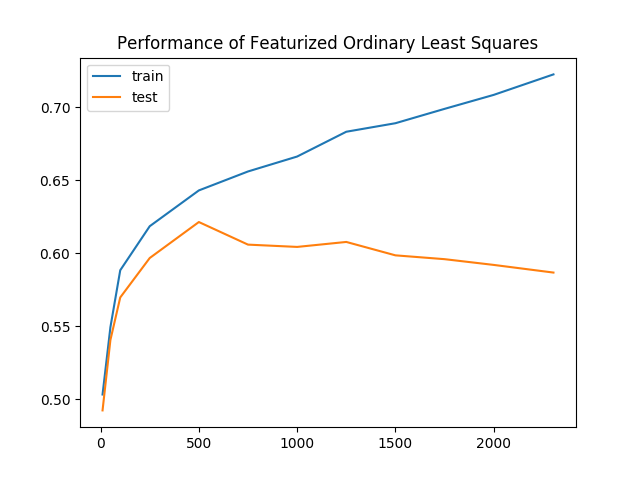As we keep more of our data, the gap between the training and validation accuracies increases as well. This is clear evidence of overfitting, where our model is learning representations that are no longer generalizable to all data. To combat overfitting, we’ll regularize our model by penalizing complex models.

We amend our ordinary least-squares objective function with a regularization term, giving us a new objective. Our new objective function is called ridge regression and it looks like this:

``````min_w |Aw- y|^2 + lambda |w|^2
``````

In this equation, `lambda` is a tunable hyperparameter. Plug `lambda = 0` into the equation and ridge regression becomes least-squares. Plug `lambda = infinity` into the equation, and you’ll find the best `w` must now be zero, as any non-zero `w` incurs infinite loss. As it turns out, this objective yields a closed-form solution as well:

``````w^* = (A^TA + lambda I)^{-1}A^Ty
``````

Still using the featurized samples, retrain and reevaluate the model once more.

Open `step_6_ls_simple.py` again in your editor:

``````nano step_6_ls_simple.py

``````

This time, increase the dimensionality of the new feature space to `d=1000​`. Change the value of `d` from `100` to `1000` as shown in the following code block:

step_6_ls_simple.py
``````...
d = 1000
W = np.random.normal(size=(X_train.shape, d))
...
``````

Then apply ridge regression using a regularization of `lambda = 10^{10}`. Replace the line defining `w` with the following two lines:

step_6_ls_simple.py
``````...
# train model
I = np.eye(A_train.shape)
w = np.linalg.inv(A_train.T.dot(A_train) + 1e10 * I).dot(A_train.T.dot(Y_oh_train))
``````

Then locate this block:

step_6_ls_simple.py
``````...
ols_train_accuracy = evaluate(A_train, Y_train, w)
print('(ols) Train Accuracy:', ols_train_accuracy)
ols_test_accuracy = evaluate(A_test, Y_test, w)
print('(ols) Test Accuracy:', ols_test_accuracy)
``````

Replace it with the following:

step_6_ls_simple.py
``````...

print('(ridge) Train Accuracy:', evaluate(A_train, Y_train, w))
print('(ridge) Test Accuracy:', evaluate(A_test, Y_test, w))
``````

The completed script should look like this:

step_6_ls_simple.py
``````"""Train emotion classifier using least squares."""

import numpy as np

def evaluate(A, Y, w):
Yhat = np.argmax(A.dot(w), axis=1)
return np.sum(Yhat == Y) / Y.shape

def main():
X_train, Y_train = data['X'], data['Y']

X_test, Y_test = data['X'], data['Y']

# one-hot labels
I = np.eye(6)
Y_oh_train, Y_oh_test = I[Y_train], I[Y_test]
d = 1000
W = np.random.normal(size=(X_train.shape, d))
# select first 100 dimensions
A_train, A_test = X_train.dot(W), X_test.dot(W)

# train model
I = np.eye(A_train.shape)
w = np.linalg.inv(A_train.T.dot(A_train) + 1e10 * I).dot(A_train.T.dot(Y_oh_train))

# evaluate model
print('(ridge) Train Accuracy:', evaluate(A_train, Y_train, w))
print('(ridge) Test Accuracy:', evaluate(A_test, Y_test, w))

if __name__ == '__main__':
main()
``````

Save the file, exit your editor, and run the script:

``````python step_6_ls_simple.py

``````

You’ll see the following output:

``````Output(ridge) Train Accuracy: 0.651173462698
(ridge) Test Accuracy: 0.622181436812
``````

There’s an additional improvement of 0.4% in validation accuracy to 62.2%, as train accuracy drops to 65.1%. Once again reevaluating across a number of different `d`, we see a smaller gap between training and validation accuracies for ridge regression. In other words, ridge regression was subject to less overfitting.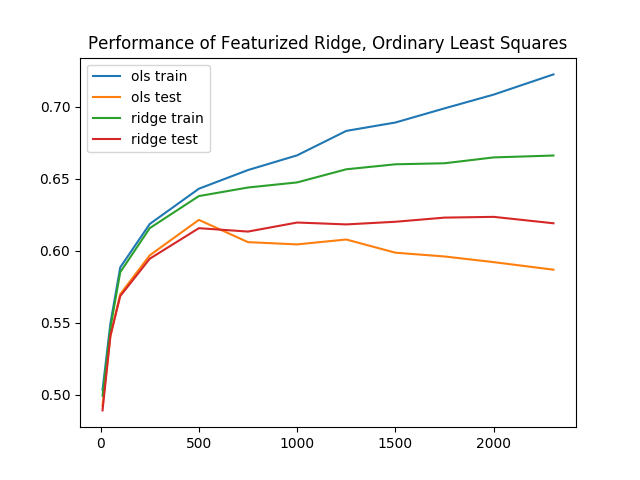Baseline performance for least squares, with these extra enhancements, performs reasonably well. The training and inference times, all together, take no more than 20 seconds for even the best results. In the next section, you’ll explore even more complex models.

## Step 7 — Building the Face-Emotion Classifier Using a Convolutional Neural Network in PyTorch

In this section, you’ll build a second emotion classifier using neural networks instead of least squares. Again, our goal is to produce a model that accepts faces as input and outputs an emotion. Eventually, this classifier will then determine which dog mask to apply.

For a brief neural network visualization and introduction, see the article Understanding Neural Networks. Here, we will use a deep-learning library called PyTorch. There are a number of deep-learning libraries in widespread use, and each has various pros and cons. PyTorch is a particularly good place to start. To impliment this neural network classifier, we again take three steps, as we did with the least-squares classifier:

1. Preprocess the data: Apply one-hot encoding and then apply PyTorch abstractions.
2. Specify and train the model: Set up a neural network using PyTorch layers. Define optimization hyperparameters and run stochastic gradient descent.
3. Run a prediction using the model: Evaluate the neural network.

Create a new file, named `step_7_fer_simple.py`

``````nano step_7_fer_simple.py

``````

Import the necessary utilities and create a Python class that will hold your data. For data processing here, you will create the train and test datasets. To do these, implement PyTorch’s `Dataset` interface, which lets you load and use PyTorch’s built-in data pipeline for the face-emotion recognition dataset:

step_7_fer_simple.py
``````from torch.utils.data import Dataset
import torch.nn as nn
import torch.nn.functional as F
import torch.optim as optim
import numpy as np
import torch
import cv2
import argparse

class Fer2013Dataset(Dataset):
"""Face Emotion Recognition dataset.

and Aaron Courville in 2013.

Each sample is 1 x 1 x 48 x 48, and each label is a scalar.
"""
pass
``````

Delete the `pass` placeholder in the `Fer2013Dataset` class. In its place, add a function that will initialize our data holder:

step_7_fer_simple.py
``````    def __init__(self, path: str):
"""
Args:
path: Path to `.np` file containing sample nxd and label nx1
"""
self._samples = data['X']
self._labels = data['Y']
self._samples = self._samples.reshape((-1, 1, 48, 48))

self.X = Variable(torch.from_numpy(self._samples)).float()
self.Y = Variable(torch.from_numpy(self._labels)).float()
...
``````

This function starts by loading the samples and labels. Then it wraps the data in PyTorch data structures.

Directly after the `__init__` function, add a `__len__` function, as this is needed to implement the `Dataset` interface PyTorch expects:

step_7_fer_simple.py
``````...
def __len__(self):
return len(self._labels)
``````

Finally, add a `__getitem__` method, which returns a dictionary containing the sample and the label:

step_7_fer_simple.py
``````    def __getitem__(self, idx):
return {'image': self._samples[idx], 'label': self._labels[idx]}
``````

Double-check that your file looks like the following:

step_7_fer_simple.py
``````from torch.utils.data import Dataset
import torch.nn as nn
import torch.nn.functional as F
import torch.optim as optim
import numpy as np
import torch
import cv2
import argparse

class Fer2013Dataset(Dataset):
"""Face Emotion Recognition dataset.
and Aaron Courville in 2013.
Each sample is 1 x 1 x 48 x 48, and each label is a scalar.
"""

def __init__(self, path: str):
"""
Args:
path: Path to `.np` file containing sample nxd and label nx1
"""
self._samples = data['X']
self._labels = data['Y']
self._samples = self._samples.reshape((-1, 1, 48, 48))

self.X = Variable(torch.from_numpy(self._samples)).float()
self.Y = Variable(torch.from_numpy(self._labels)).float()

def __len__(self):
return len(self._labels)

def __getitem__(self, idx):
return {'image': self._samples[idx], 'label': self._labels[idx]}
``````

Next, load the `Fer2013Dataset` dataset. Add the following code to the end of your file after the `Fer2013Dataset` class:

step_7_fer_simple.py
``````trainset = Fer2013Dataset('data/fer2013_train.npz')

testset = Fer2013Dataset('data/fer2013_test.npz')
``````

This code initializes the dataset using the `Fer2013Dataset` class you created. Then for the train and validation sets, it wraps the dataset in a `DataLoader`. This translates the dataset into an iterable to use later.

As a sanity check, verify that the dataset utilities are functioning. Create a sample dataset loader using `DataLoader` and print the first element of that loader. Add the following to the end of your file:

step_7_fer_simple.py
``````if __name__ == '__main__':
``````

Verify that your completed script looks like this:

step_7_fer_simple.py
``````from torch.utils.data import Dataset
import torch.nn as nn
import torch.nn.functional as F
import torch.optim as optim
import numpy as np
import torch
import cv2
import argparse

class Fer2013Dataset(Dataset):
"""Face Emotion Recognition dataset.
and Aaron Courville in 2013.
Each sample is 1 x 1 x 48 x 48, and each label is a scalar.
"""

def __init__(self, path: str):
"""
Args:
path: Path to `.np` file containing sample nxd and label nx1
"""
self._samples = data['X']
self._labels = data['Y']
self._samples = self._samples.reshape((-1, 1, 48, 48))

self.X = Variable(torch.from_numpy(self._samples)).float()
self.Y = Variable(torch.from_numpy(self._labels)).float()

def __len__(self):
return len(self._labels)

def __getitem__(self, idx):
return {'image': self._samples[idx], 'label': self._labels[idx]}

trainset = Fer2013Dataset('data/fer2013_train.npz')

testset = Fer2013Dataset('data/fer2013_test.npz')

if __name__ == '__main__':
``````

Exit your editor and run the script.

``````python step_7_fer_simple.py

``````

This outputs the following pair of tensors. Our data pipeline outputs two samples and two labels. This indicates that our data pipeline is up and ready to go:

``````Output{'image':
(0 ,0 ,.,.) =
24   32   36  ...   173  172  173
25   34   29  ...   173  172  173
26   29   25  ...   172  172  174
...         ⋱        ...
159  185  157  ...   157  156  153
136  157  187  ...   152  152  150
145  130  161  ...   142  143  142
⋮

(1 ,0 ,.,.) =
20   17   19  ...   187  176  162
22   17   17  ...   195  180  171
17   17   18  ...   203  193  175
...         ⋱        ...
1    1    1  ...   106  115  119
2    2    1  ...   103  111  119
2    2    2  ...    99  107  118
[torch.LongTensor of size 2x1x48x48]
, 'label':
1
1
[torch.LongTensor of size 2]
}
``````

Now that you’ve verified that the data pipeline works, return to `step_7_fer_simple.py` to add the neural network and optimizer. Open `step_7_fer_simple.py`.

``````nano step_7_fer_simple.py

``````

First, delete the last three lines you added in the previous iteration:

step_7_fer_simple.py
``````# Delete all three lines
if __name__ == '__main__':
``````

In their place, define a PyTorch neural network that includes three convolutional layers, followed by three fully connected layers. Add this to the end of your existing script:

step_7_fer_simple.py
``````class Net(nn.Module):
def __init__(self):
super(Net, self).__init__()
self.conv1 = nn.Conv2d(1, 6, 5)
self.pool = nn.MaxPool2d(2, 2)
self.conv2 = nn.Conv2d(6, 6, 3)
self.conv3 = nn.Conv2d(6, 16, 3)
self.fc1 = nn.Linear(16 * 4 * 4, 120)
self.fc2 = nn.Linear(120, 48)
self.fc3 = nn.Linear(48, 3)

def forward(self, x):
x = self.pool(F.relu(self.conv1(x)))
x = self.pool(F.relu(self.conv2(x)))
x = self.pool(F.relu(self.conv3(x)))
x = x.view(-1, 16 * 4 * 4)
x = F.relu(self.fc1(x))
x = F.relu(self.fc2(x))
x = self.fc3(x)
return x
``````

Now initialize the neural network, define a loss function, and define optimization hyperparameters by adding the following code to the end of the script:

step_7_fer_simple.py
``````net = Net().float()
criterion = nn.CrossEntropyLoss()
optimizer = optim.SGD(net.parameters(), lr=0.001, momentum=0.9)
``````

We’ll train for two epochs. For now, we define an epoch to be an iteration of training where every training sample has been used exactly once.

First, extract `image` and `label` from the dataset loader and then wrap each in a PyTorch `Variable`. Second, run the forward pass and then backpropagate through the loss and neural network. Add the following code to the end of your script to do that:

step_7_fer_simple.py
``````for epoch in range(2):  # loop over the dataset multiple times

running_loss = 0.0
for i, data in enumerate(trainloader, 0):
inputs = Variable(data['image'].float())
labels = Variable(data['label'].long())

# forward + backward + optimize
outputs = net(inputs)
loss = criterion(outputs, labels)
loss.backward()
optimizer.step()

# print statistics
running_loss += loss.data
if i % 100 == 0:
print('[%d, %5d] loss: %.3f' % (epoch, i, running_loss / (i + 1)))
``````

Your script should now look like this:

step_7_fer_simple.py
``````from torch.utils.data import Dataset
import torch.nn as nn
import torch.nn.functional as F
import torch.optim as optim
import numpy as np
import torch
import cv2
import argparse

class Fer2013Dataset(Dataset):
"""Face Emotion Recognition dataset.

and Aaron Courville in 2013.

Each sample is 1 x 1 x 48 x 48, and each label is a scalar.
"""
def __init__(self, path: str):
"""
Args:
path: Path to `.np` file containing sample nxd and label nx1
"""
self._samples = data['X']
self._labels = data['Y']
self._samples = self._samples.reshape((-1, 1, 48, 48))

self.X = Variable(torch.from_numpy(self._samples)).float()
self.Y = Variable(torch.from_numpy(self._labels)).float()

def __len__(self):
return len(self._labels)

def __getitem__(self, idx):
return {'image': self._samples[idx], 'label': self._labels[idx]}

trainset = Fer2013Dataset('data/fer2013_train.npz')

testset = Fer2013Dataset('data/fer2013_test.npz')

class Net(nn.Module):
def __init__(self):
super(Net, self).__init__()
self.conv1 = nn.Conv2d(1, 6, 5)
self.pool = nn.MaxPool2d(2, 2)
self.conv2 = nn.Conv2d(6, 6, 3)
self.conv3 = nn.Conv2d(6, 16, 3)
self.fc1 = nn.Linear(16 * 4 * 4, 120)
self.fc2 = nn.Linear(120, 48)
self.fc3 = nn.Linear(48, 3)

def forward(self, x):
x = self.pool(F.relu(self.conv1(x)))
x = self.pool(F.relu(self.conv2(x)))
x = self.pool(F.relu(self.conv3(x)))
x = x.view(-1, 16 * 4 * 4)
x = F.relu(self.fc1(x))
x = F.relu(self.fc2(x))
x = self.fc3(x)
return x

net = Net().float()
criterion = nn.CrossEntropyLoss()
optimizer = optim.SGD(net.parameters(), lr=0.001, momentum=0.9)

for epoch in range(2):  # loop over the dataset multiple times

running_loss = 0.0
for i, data in enumerate(trainloader, 0):
inputs = Variable(data['image'].float())
labels = Variable(data['label'].long())

# forward + backward + optimize
outputs = net(inputs)
loss = criterion(outputs, labels)
loss.backward()
optimizer.step()

# print statistics
running_loss += loss.data
if i % 100 == 0:
print('[%d, %5d] loss: %.3f' % (epoch, i, running_loss / (i + 1)))
``````

Save the file and exit the editor once you’ve verified your code. Then, launch this proof-of-concept training:

``````python step_7_fer_simple.py

``````

You’ll see output similar to the following as the neural network trains:

``````Output[0,     0] loss: 1.094
[0,   100] loss: 1.049
[0,   200] loss: 1.009
[0,   300] loss: 0.963
[0,   400] loss: 0.935
[1,     0] loss: 0.760
[1,   100] loss: 0.768
[1,   200] loss: 0.775
[1,   300] loss: 0.776
[1,   400] loss: 0.767
``````

You can then augment this script using a number of other PyTorch utilities to save and load models, output training and validation accuracies, fine-tune a learning-rate schedule, etc. After training for 20 epochs with a learning rate of 0.01 and momentum of 0.9, our neural network attains a 87.9% train accuracy and a 75.5% validation accuracy, a further 6.8% improvement over the most successful least-squares approach thus far at 66.6%. We’ll include these additional bells and whistles in a new script.

Create a new file to hold the final face emotion detector which your live camera feed will use. This script contains the code above along with a command-line interface and an easy-to-import version of our code that will be used later. Additionally, it contains the hyperparameters tuned in advance, for a model with higher accuracy.

``````nano step_7_fer.py

``````

Start with the following imports. This matches our previous file but additionally includes OpenCV as `import cv2.`

step_7_fer.py
``````from torch.utils.data import Dataset
import torch.nn as nn
import torch.nn.functional as F
import torch.optim as optim
import numpy as np
import torch
import cv2
import argparse
``````

Directly beneath these imports, reuse your code from `step_7_fer_simple.py` to define the neural network:

step_7_fer.py
``````class Net(nn.Module):
def __init__(self):
super(Net, self).__init__()
self.conv1 = nn.Conv2d(1, 6, 5)
self.pool = nn.MaxPool2d(2, 2)
self.conv2 = nn.Conv2d(6, 6, 3)
self.conv3 = nn.Conv2d(6, 16, 3)
self.fc1 = nn.Linear(16 * 4 * 4, 120)
self.fc2 = nn.Linear(120, 48)
self.fc3 = nn.Linear(48, 3)

def forward(self, x):
x = self.pool(F.relu(self.conv1(x)))
x = self.pool(F.relu(self.conv2(x)))
x = self.pool(F.relu(self.conv3(x)))
x = x.view(-1, 16 * 4 * 4)
x = F.relu(self.fc1(x))
x = F.relu(self.fc2(x))
x = self.fc3(x)
return x
``````

Again, reuse the code for the Face Emotion Recognition dataset from `step_7_fer_simple.py` and add it to this file:

step_7_fer.py
``````class Fer2013Dataset(Dataset):
"""Face Emotion Recognition dataset.
and Aaron Courville in 2013.
Each sample is 1 x 1 x 48 x 48, and each label is a scalar.
"""

def __init__(self, path: str):
"""
Args:
path: Path to `.np` file containing sample nxd and label nx1
"""
self._samples = data['X']
self._labels = data['Y']
self._samples = self._samples.reshape((-1, 1, 48, 48))

self.X = Variable(torch.from_numpy(self._samples)).float()
self.Y = Variable(torch.from_numpy(self._labels)).float()

def __len__(self):
return len(self._labels)

def __getitem__(self, idx):
return {'image': self._samples[idx], 'label': self._labels[idx]}
``````

Next, define a few utilities to evaluate the neural network’s performance. First, add an `evaluate` function which compares the neural network’s predicted emotion to the true emotion for a single image:

step_7_fer.py
``````def evaluate(outputs: Variable, labels: Variable, normalized: bool=True) -> float:
"""Evaluate neural network outputs against non-one-hotted labels."""
Y = labels.data.numpy()
Yhat = np.argmax(outputs.data.numpy(), axis=1)
denom = Y.shape if normalized else 1
return float(np.sum(Yhat == Y) / denom)
``````

Then add a function called `batch_evaluate` which applies the first function to all images:

step_7_fer.py
``````def batch_evaluate(net: Net, dataset: Dataset, batch_size: int=500) -> float:
"""Evaluate neural network in batches, if dataset is too large."""
score = 0.0
n = dataset.X.shape
for i in range(0, n, batch_size):
x = dataset.X[i: i + batch_size]
y = dataset.Y[i: i + batch_size]
score += evaluate(net(x), y, False)
return score / n
``````

Now, define a function called `get_image_to_emotion_predictor` that takes in an image and outputs a predicted emotion, using a pretrained model:

step_7_fer.py
``````def get_image_to_emotion_predictor(model_path='assets/model_best.pth'):
"""Returns predictor, from image to emotion index."""
net = Net().float()

def predictor(image: np.array):
"""Translates images into emotion indices."""
if image.shape > 1:
image = cv2.cvtColor(image, cv2.COLOR_BGR2GRAY)
frame = cv2.resize(image, (48, 48)).reshape((1, 1, 48, 48))
X = Variable(torch.from_numpy(frame)).float()
return np.argmax(net(X).data.numpy(), axis=1)
return predictor
``````

Finally, add the following code to define the `main` function to leverage the other utilities:

step_7_fer.py
``````def main():
trainset = Fer2013Dataset('data/fer2013_train.npz')
testset = Fer2013Dataset('data/fer2013_test.npz')
net = Net().float()

train_acc = batch_evaluate(net, trainset, batch_size=500)
print('Training accuracy: %.3f' % train_acc)
test_acc = batch_evaluate(net, testset, batch_size=500)
print('Validation accuracy: %.3f' % test_acc)

if __name__ == '__main__':
main()
``````

This loads a pretrained neural network and evaluates its performance on the provided Face Emotion Recognition dataset. Specifically, the script outputs accuracy on the images we used for training, as well as a separate set of images we put aside for testing purposes.

Double-check that your file matches the following:

step_7_fer.py
``````from torch.utils.data import Dataset
import torch.nn as nn
import torch.nn.functional as F
import torch.optim as optim
import numpy as np
import torch
import cv2
import argparse

class Net(nn.Module):
def __init__(self):
super(Net, self).__init__()
self.conv1 = nn.Conv2d(1, 6, 5)
self.pool = nn.MaxPool2d(2, 2)
self.conv2 = nn.Conv2d(6, 6, 3)
self.conv3 = nn.Conv2d(6, 16, 3)
self.fc1 = nn.Linear(16 * 4 * 4, 120)
self.fc2 = nn.Linear(120, 48)
self.fc3 = nn.Linear(48, 3)

def forward(self, x):
x = self.pool(F.relu(self.conv1(x)))
x = self.pool(F.relu(self.conv2(x)))
x = self.pool(F.relu(self.conv3(x)))
x = x.view(-1, 16 * 4 * 4)
x = F.relu(self.fc1(x))
x = F.relu(self.fc2(x))
x = self.fc3(x)
return x

class Fer2013Dataset(Dataset):
"""Face Emotion Recognition dataset.
and Aaron Courville in 2013.
Each sample is 1 x 1 x 48 x 48, and each label is a scalar.
"""

def __init__(self, path: str):
"""
Args:
path: Path to `.np` file containing sample nxd and label nx1
"""
self._samples = data['X']
self._labels = data['Y']
self._samples = self._samples.reshape((-1, 1, 48, 48))

self.X = Variable(torch.from_numpy(self._samples)).float()
self.Y = Variable(torch.from_numpy(self._labels)).float()

def __len__(self):
return len(self._labels)

def __getitem__(self, idx):
return {'image': self._samples[idx], 'label': self._labels[idx]}

def evaluate(outputs: Variable, labels: Variable, normalized: bool=True) -> float:
"""Evaluate neural network outputs against non-one-hotted labels."""
Y = labels.data.numpy()
Yhat = np.argmax(outputs.data.numpy(), axis=1)
denom = Y.shape if normalized else 1
return float(np.sum(Yhat == Y) / denom)

def batch_evaluate(net: Net, dataset: Dataset, batch_size: int=500) -> float:
"""Evaluate neural network in batches, if dataset is too large."""
score = 0.0
n = dataset.X.shape
for i in range(0, n, batch_size):
x = dataset.X[i: i + batch_size]
y = dataset.Y[i: i + batch_size]
score += evaluate(net(x), y, False)
return score / n

def get_image_to_emotion_predictor(model_path='assets/model_best.pth'):
"""Returns predictor, from image to emotion index."""
net = Net().float()

def predictor(image: np.array):
"""Translates images into emotion indices."""
if image.shape > 1:
image = cv2.cvtColor(image, cv2.COLOR_BGR2GRAY)
frame = cv2.resize(image, (48, 48)).reshape((1, 1, 48, 48))
X = Variable(torch.from_numpy(frame)).float()
return np.argmax(net(X).data.numpy(), axis=1)
return predictor

def main():
trainset = Fer2013Dataset('data/fer2013_train.npz')
testset = Fer2013Dataset('data/fer2013_test.npz')
net = Net().float()

train_acc = batch_evaluate(net, trainset, batch_size=500)
print('Training accuracy: %.3f' % train_acc)
test_acc = batch_evaluate(net, testset, batch_size=500)
print('Validation accuracy: %.3f' % test_acc)

if __name__ == '__main__':
main(
``````

Save the file and exit your editor.

As before, with the face detector, download pre-trained model parameters and save them to your `assets` folder with the following command:

``````wget -O assets/model_best.pth https://github.com/alvinwan/emotion-based-dog-filter/raw/master/src/assets/model_best.pth

``````

Run the script to use and evaluate the pre-trained model:

``````python step_7_fer.py

``````

This will output the following:

``````OutputTraining accuracy: 0.879
Validation accuracy: 0.755
``````

At this point, you’ve built a pretty accurate face-emotion classifier. In essence, our model can correctly disambiguate between faces that are happy, sad, and surprised eight out of ten times. This is a reasonably good model, so you can now move on to using this face-emotion classifier to determine which dog mask to apply to faces.

## Step 8 — Finishing the Emotion-Based Dog Filter

Before integrating our brand-new face-emotion classifier, we will need animal masks to pick from. We’ll use a Dalmation mask and a Sheepdog mask: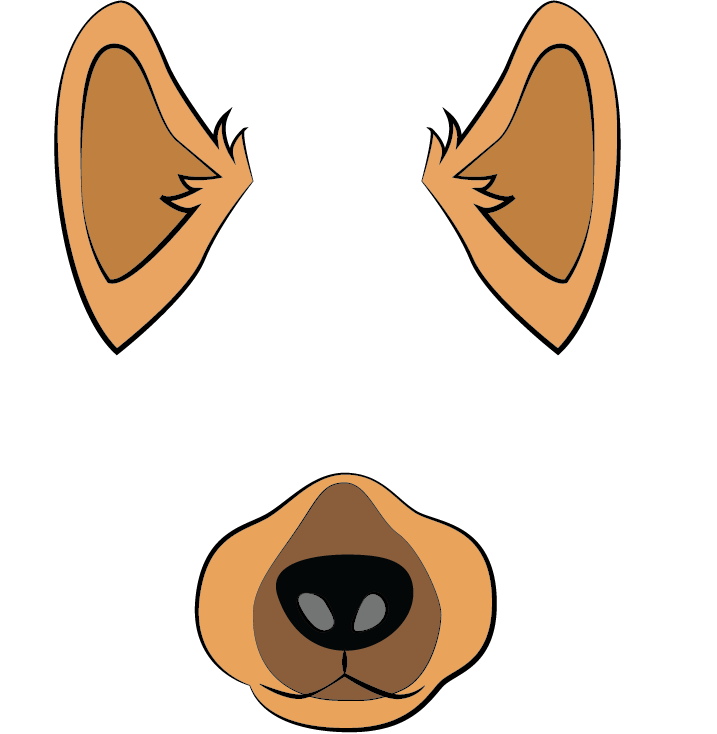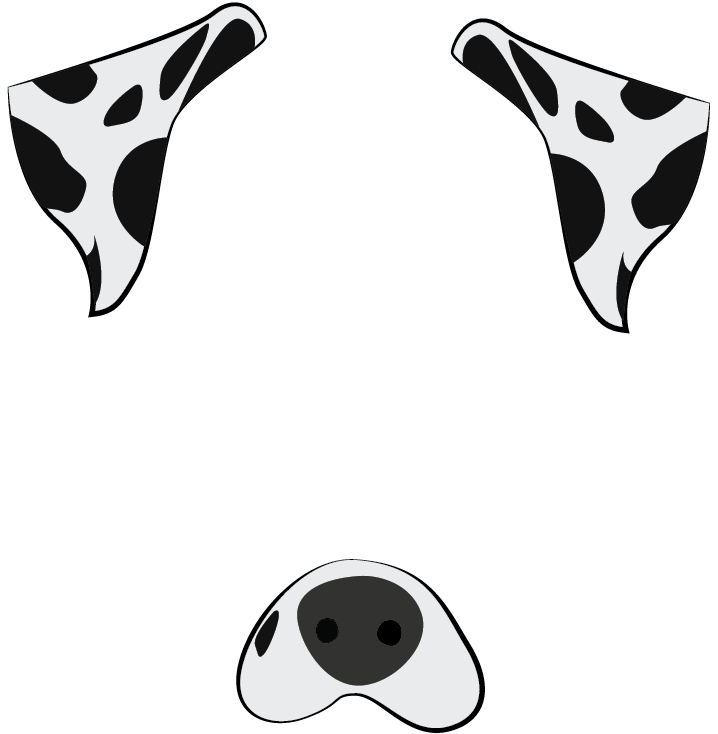Execute these commands to download both masks to your `assets` folder:

``````wget -O assets/dalmation.png https://assets.digitalocean.com/articles/python3_dogfilter/E9ax7PI.png  # dalmation
wget -O assets/sheepdog.png https://assets.digitalocean.com/articles/python3_dogfilter/HveFdkg.png  # sheepdog

``````

Now let’s use the masks in our filter. Start by duplicating the `step_4_dog_mask.py` file:

``````cp step_4_dog_mask.py step_8_dog_emotion_mask.py

``````

Open the new Python script.

``````nano step_8_dog_emotion_mask.py

``````

Insert a new line at the top of the script to import the emotion predictor:

``````from step_7_fer import get_image_to_emotion_predictor
...
``````

Then, in the `main()` function, locate this line:

``````    mask = cv2.imread('assets/dog.png')
``````

Replace it with the following to load the new masks and aggregate all masks into a tuple:

``````    mask0 = cv2.imread('assets/dog.png')
``````

Add a line break, and then add this code to create the emotion predictor.

``````
# get emotion predictor
predictor = get_image_to_emotion_predictor()
``````

Your `main` function should now match the following:

``````def main():
cap = cv2.VideoCapture(0)

``````